# How to glue derived categories

## Abstract

We give an overview of existing enhancement techniques for derived and trianguated categories based on the notion of a stable model category, and show how it can be applied to the problem of gluing triangulated categories. The article is mostly expository, but we do prove some new results concerning existence of model structures.

## Introduction and historical overview

The basic question addressed in this paper is formulated in the title, and it looks extremely old-fashioned: while in the early 1990-ies, this would have been a hot research topic, by now so many solutions are available in the literature that the question looks completely closed. To explain why in our view the question is still relevant, let us start with a brief historical overview.

### Derived categories

From its very beginning [10, 20], homological algebra was based on the notion of a right and left-derived functor. For right-derived functors, one starts with a left-exact functor $$F:\mathcal {C}\rightarrow \mathcal {E}$$ between abelian categories $$\mathcal {C}$$, $$\mathcal {E}$$. To construct the right-derived functors $$R^\cdot F$$, one replaces an object $$c \in \mathcal {C}$$ by an appropriate (for example, injective) resolution, applies F, and takes cohomology. For left-derived functors, the definition is dual. By a general theorem, the result of the procedure does not depend on the choice of a resolution.

The formalism of derived categories that appeared in  internalizes this independence: to define the derived category $${\mathcal {D}}(\mathcal {C})$$, one takes the category $$C_\cdot (\mathcal {C})$$ of chain complexes of objects in $$\mathcal {C}$$, and formally inverts quasiisomorphisms. Since two resolutions of the same object are quasiisomorphic, they give the same object in $${\mathcal {D}}(\mathcal {C})$$. Therefore $${\mathcal {D}}(\mathcal {C})$$ is the natural domain of definition for derived functors.

The procedure of formally inverting a class of maps in a category is known as localization, and it is rather non-trivial. For $$C_\cdot (\mathcal {C})$$, one does it in two steps. First, one considers the category $$H(\mathcal {C})$$ whose objects are chain complexes in $$\mathcal {C}$$, and whose maps are chain-homotopy equivalence classes of maps in $$C_\cdot (\mathcal {C})$$. Then one localizes $$H(\mathcal {C})$$ to obtain $${\mathcal {D}}(\mathcal {C})$$. For the second step, one uses an additional structure carried by $$H(\mathcal {C})$$ and $${\mathcal {D}}(\mathcal {C})$$—that of a triangulated category. This notion also appeared in , following an earlier version in . The crucial difference is the so-called octahedron axiom missing in  that allows one to prove the Verdier Localization Theorem: given a full triangulated subcategory $${\mathcal {D}}' \subset {\mathcal {D}}$$ in a triangulated category $${\mathcal {D}}$$ satisfying some mild conditions, one can construct a triangulated “quotient category” $${\mathcal {D}}/{\mathcal {D}}'$$ that is the localization of $${\mathcal {D}}$$ with respect to all maps whose cone lies in $${\mathcal {D}}'$$. To obtain $${\mathcal {D}}(\mathcal {C})$$, one takes $${\mathcal {D}}=H(\mathcal {C})$$, and lets $${\mathcal {D}}'$$ be the full subcategory spanned by acyclic complexes.

### Triangulated categories

In the decades that passed since , derived and triangulated categories have enjoyed a lot of success in several areas of mathematics. Already by 1980-ies, they have become ubiquitous at least in algebraic geometry and representation theory. For derived categories, this is not surprising, since many fundamental results such as the Grothendieck-Serre Duality cannot even be formulated without using them. As for the triangulated structure, two reasons for its success come to mind:

• Ease of construction. Any condition on objects in a triangulated category $${\mathcal {D}}$$ closed under taking cones defines a full triangulated subcategory $${\mathcal {D}}' \subset {\mathcal {D}}$$; if needed, one can then take the quotient $${\mathcal {D}}/{\mathcal {D}}'$$, find another full subcategory inside it, and so on.

• Ease of comparison. For example, assume given triangulated categories $${\mathcal {D}}$$, $${\mathcal {D}}'$$ with generators $$E \in {\mathcal {D}}$$, $$E' \in {\mathcal {D}}'$$, and a triangulated functor $$F:{\mathcal {D}}' \rightarrow {\mathcal {D}}$$ such that $$F(E')=E$$. Then to check that F is an equivalence, it suffices to prove that the induced map $$F:{\text {Hom}}(E',E'[n]) \rightarrow {\text {Hom}}(E,E[n])$$ is an isomorphism for any integer n.

The construction methods described above easily take one beyond derived categories, and this is often indispensable—for example, the category of étale sheaves of $$\mathbb {Q}_l$$-vector spaces is not the derived category of anything at all. On the other hand, the same triangulated category $${\mathcal {D}}$$ can appear as the derived category of different abelian ones (if needed, these can be located inside $${\mathcal {D}}$$ by the machinery of t-structures of ). All this and more is discussed at length in , the first textbook dedicated specifically to derived categories, and a great snapshot of the state-of-the-art in the subject at the time of its publication.

However, it became clear very soon that the notion of a triangulated category has grave deficiencies: several important constructions that work for abelian categories stop working in the triangulated setting. For example, if we have a left-exact functor $$F:\mathcal {C}\rightarrow \mathcal {C}'$$ between abelian categories, then the category $$\widetilde{\mathcal {C}}$$ of triples $$\langle c,c',f \rangle$$, $$c \in \mathcal {C}$$, $$c' \in \mathcal {C}'$$, $$f:c' \rightarrow F(c)$$ is abelian (this is known as gluing). The corresponding statement for triangulated categories is not true. Worse than that, there is no way to recover $${\mathcal {D}}(\widetilde{\mathcal {C}})$$ from $${\mathcal {D}}(\mathcal {C})$$, $${\mathcal {D}}(\mathcal {C}')$$ and the derived functor $$R^\cdot F:{\mathcal {D}}(\mathcal {C}) \rightarrow {\mathcal {D}}(\mathcal {C}')$$.

More generally, the notion of a family of categories parametrized by a small category I is conveniently formalized as a fibration $$\mathcal {C}\rightarrow I$$ in the sense of  (a Grothendieck fibration). Then if the fibers $$\mathcal {C}_i$$, $$i \in I$$ of the fibration are abelian, and the transition functors are left-exact, the category $${\text {Sec}}(\mathcal {C})$$ of sections $$I \rightarrow \mathcal {C}$$ of the fibration $$\mathcal {C}\rightarrow I$$ is abelian. For triangulated categories, the statement is wrong, and moreover, one cannot recover the derived category $${\mathcal {D}}({\text {Sec}}(\mathcal {C}))$$ from the family of the derived categories $${\mathcal {D}}(\mathcal {C}_i)$$, $$i \in I$$. This happens even when the family is the constant family $$\mathcal {C}= \mathcal {C}_0 \times I$$, so that $${\text {Sec}}(\mathcal {C})$$ is the category $$\mathcal {C}_0^I$$ of functors from I to an abelian category $$\mathcal {C}_0$$: one cannot recover $${\mathcal {D}}(\mathcal {C}_0^I)$$ from I and $${\mathcal {D}}(\mathcal {C}_0)$$.

One can say that the structure of a triangulated category captures some of the natural structure possessed by a derived category $${\mathcal {D}}(\mathcal {C})$$ but not all of it; a satisfactory notion would be a triangulated category “with enhancement”.

Unfortunately, finding out what this “enhancement” might be, exactly, turned out to be an extremely difficult problem.

### Enhancements

Historically, the first notion of an enhancement was suggested by Grothendieck , based on his idea of a derivator. This is very pleasing conceptually but has technical problems; although it has been vigorously developed, it is even now perhaps not ready for practical use.

A more practical alternative is the so-called DG enhancement. For details on this, we refer the reader to  or to a comprehensive recent overview in [38, Section 2]. Here is the main idea. In the derived category case, one observes for any two objects $$c,c' \in C_\cdot (\mathcal {C})$$, we have a whole complex of morphisms $${\text {Hom}}^\cdot (c,c')$$. Maps in $$H(\mathcal {C})$$ correspond to degree-0 cohomology classes of this complex. One axiomatizes the situation by introducing a notion of a DG category $$\mathcal {C}_\cdot$$ that by definition has a complex $$C_\cdot (c,c')$$ of morphisms for any two objects. Taking degree-0 cohomology of these complexes, we obtain a category $$H^0(\mathcal {C}_\cdot )$$. One distinguishes a class of pretriangulated DG categories $$\mathcal {C}_\cdot$$ for which $$H^0(\mathcal {C}_\cdot )$$ has a natural triangulated structure. One says that a DG functor $$F:\mathcal {C}_\cdot \rightarrow \mathcal {C}_\cdot '$$ is a quasi-equivalence if $$F:\mathcal {C}_\cdot (c,c') \rightarrow \mathcal {C}'_\cdot (F(c),F(c')$$ is a quasiisomorphism for any $$c,c' \in \mathcal {C}$$, and the induced functor $$H^0(F):H^0(\mathcal {C}_\cdot ) \rightarrow H^0(\mathcal {C}'_\cdot )$$ is an equivalence. Then a DG enhancement of a triangulated category $${\mathcal {D}}$$ is a pretriangulated DG category $${\mathcal {D}}_\cdot$$ equipped with an equivalence $$H^0({\mathcal {D}}_\cdot ) \cong {\mathcal {D}}$$, considered up to a quasiequivalence.

At a first glance, this approach feels like a throwback to earlier times when one had to choose resolutions, with the only difference that now we choose all resolutions at the very beginning. Independence of this choice is now encoded in the fact that we consider DG categories up to a quasiequivalence. This is again a localization problem: we have the category of DG categories, and we want to invert quasiequivalences between them.

Since the category of DG categories is not additive, this localization problem requires new methods. Fortunately, these methods do exist, in the form of model structures and model categories introduced by Quillen .

Model categories were somewhat neglected for a while, but the subject has experienced a real resurgence starting approximately from the book , and has been very active since then. In particular, a good model structure on the category of DG categories has been constructed in , and the corresponding localized category $$\mathsf{dgcat}$$ has been studied extensively in . This gives precise meaning to the phrase “DG category up to a quasiequivalence”, and makes DG enhancements a workable tool.

It does have its drawbacks, though, and here are two of them.

1. (i)

It only work fors “algebraic” triangulated categories in the sense of ; there are many interesting categories that come from algebraic topology, and these are known not to admit DG enhancements (see e.g. ).

2. (ii)

A DG category is not a category—it is a category with an additional structure. Therefore we cannot package a family $$\mathcal {C}_\cdot$$ of DG categories parametrized by some I into a single category by the Grothendieck construction. Moreover, even if we forget the additional structure, the corresponding fibered category $$\mathcal {C}\rightarrow I$$ itself is not a DG category, and neither is the category of sections $${\text {Sec}}(\mathcal {C})$$. In particular, the category $$\mathcal {C}^I$$ of functors from a small category I to a DG category $$\mathcal {C}$$ is not a DG category. Both $${\text {Sec}}(\mathcal {C})$$ and $$\mathcal {C}^I$$ can be made into DG categories by additional technical trickery, but the construction is unpleasant and depends on irrelevant choices.

In the context of this paper, the problem (ii) is especially unpleasant, since it precludes the existence of a simple gluing procedure for DG enhanced categories. In practice—for a recent illustration, see e.g. —one glues DG categories by using the general formalism of homotopy limits in $$\mathsf{dgcat}$$. This gives an answer but very little control over this answer.

### Recent developments

Recent resurgence of interest in the model categories is actually explained by developments in algebraic topology, where the late 1990-ies saw a tremendous push to put the foundations of the subject on a more solid ground, with remarkable achievements such as “brave new algebra” of [18, 27]. It seems that at first, the notion of a triangulated category was not considered very useful in topology. However, the situation corrected itself very soon, and many versions of enhancements appropriate in the topological context appeared in the literature.

It is certainly too early to summarize this very active area. However, it probably would not be too far off the mark to say that most recent developments focus on the notion of an $$\infty$$ -category.

As an idea, this goes back to , and one way to phrase it is the following. What localization produces naturally is not just a category, it is in fact a category enriched in homotopy types: for any two objects c, $$c'$$, we have a homotopy type $${\text {Hom}}(c,c')$$ whose $$\pi _0$$ is the set of maps in the localized category (for a precise construction of $${\text {Hom}}(c,c')$$ see ). If one wants to also keep track of $$\pi _1$$, then up to an equivalence, a homotopy type can be replaced with its Poincaré groupoid. A category enriched in groupoids is a 2-category, or rather, a (2, 1)-category (all 1-morphisms are invertible). What one would like to have, then, are notions of an “$$\infty$$-groupoid” and an “$$(\infty ,1)$$-category”—higher versions of groupoids and (2, 1)-categories that keep track of all the homotopy groups. This is what localization should produce, and this is the correct structure to consider. One can further distinguish a subclass of stable $$\infty$$-categories; these are automatically enriched in stable homotopy types, produce triangulated categories after truncation, and give the correct notion of enhancement.

This idea was discussed quite a lot in the early 1990-ies, and probably before that, but people were reluctant to try to turn it into actual mathematics. Here is a rough summary explaining why:

1. (i)

It was felt that it certainly can be done, and in several ways.

2. (ii)

All of the constructions would be very complicated and ad hoc. There is no preferred construction.

3. (iii)

This would not matter: all of the constructions will be equivalent, and moreover, “the space of all possible constructions is contractible”.

4. (iv)

Moreover, one could even turn the last statement into a theorem.

5. (v)

However, to do so, one needs to make sense of “the space of all constructions”. This is certainly possible, and there are several ways to do it, just as in (i). But then one also has (ii), (iii), (iv), (v), and the infinite loop.

In the twenty years that passed, there has been a tremendous amount of research in the area, and the feelings enumerated above have been mostly confirmed (a great overview can be found in  that gets all the way to (iv) and then to (i) of the second interation). At present, there are several constructions of $$\infty$$-categories in the literature (e.g. quasicategories of Joyal as developed by Lurie [33, 34], complete Segal spaces of Rezk , and an attempt at a more invariant treatment by Barwick and Kan ). All of them are quite heavy and/or inexplicit. All of them are equivalent, but the proofs of this are also quite heavy. Some of the crucial references on the subject such as  stay unpublished for several years, presumably because they are not in a final form. Nevertheless, the theory does exist, and when applied to triangulated categories, it does provide a notion of enhancement in the from of a stable $$\infty$$-category. Being explicitly topological in origin, this type of enhancement is certainly free from the first problem of DG enhancements mentioned above.

In a way, it is also free from the second problem, but this comes at a price. Effectively, one observes that a category is also a $$\infty$$-category, and a family $$\mathcal {C}_\cdot$$ of $$\infty$$-categories indexed by some I can be turned into a single $$\infty$$-functor $$\mathcal {C}\rightarrow I$$ by an $$\infty$$-version of the Grothendieck construction. Then to glue the family, one can again consider the sections of this functor. However, to get the correct result, these must be $$\infty$$-sections. By a general theorem, these exist and form an $$\infty$$-category, stable if so where the fibers $$\mathcal {C}_i$$, but this is all one can say for free. Getting any sort of control over the result of the procedure requires one to actually go into the definitions, and none of them are easy or constructive.

In practice, people try to use the machinery of $$\infty$$-categories as a black box, but the results are somewhat mixed—it is hard to work with a black box that contains not only proofs but also statements and definitions. It seems that the $$\infty$$-technology as presently developed is of an all-or-nothing type: either we move the whole of mathematics to the $$\infty$$-categorical setting, or we cannot use it at all. This might have been tempting, were the setting really simple or really natural, but it is neither, with a lot of pretty arbitrary choices hardcoded into its very foundations. So, while the move might happen eventually, now is clearly not the time.

It is unlikely to happen in near future either, since, specific choice of models aside, we do not understand some very basic things. Let me list two.

1. (i)

Why do we have to replace sets with simplicial sets? Chain complexes and cohomology long exact sequences emerge naturally when one considers functors exact on one side, and tries to find obstructions to exactness. The category $$\Delta$$ appears quite naturally in algebra and category theory (e.g. [37, VII.5]); the fact that it has something to do with derived functors and enhancements is just postulated. We have a pretty good idea of why simplicial sets give a good model for topological spaces, and vice versa (see e.g.  or ), so to a topologist, there is no problem; everybody else has to just take it on faith.

2. (ii)

Why do we need to replace the naive localization with the Dwyer–Kan localization? The motivation given by Dwyer and Kan is group completion: for a free monoid M, its group completion $$\Omega BM$$ is just the free group, and if M is not free, it can have higher homotopy. For a topologist, or at least for someone with some experience of topology, this is quite convincing. For everybody else, this makes no sense: why would one involve $$\Omega BM$$, a topological space, in such a purely algebraic question? Tensor product is exact on the right and not on the left, so we have to derive it; an instrinsic explanation of why we have to derive localization is not in the literature.

An approach to the subject in the spirit of  would address and resolve at least these foundational issues, and then develop the formalism from there. The existing approaches declare the issues solved by consensus. As a result, they are forcing things instead of trying to understand them, and get mired in technical intricacies of simplicial homotopy theory, cofibrantly generated model categories, small object arguments and suchlike. Within topology, this is perfectly fine, since it works, and nothing else does. However, triangulated categories are widely used outside of topology, and an attempt to impose all the idiosyncratic machinery on experts in algebraic geometry or representation theory seems hardly fair, and rather futile.

### The content of the paper

Let us now explain what we do in this paper. We certainly do not attempt to suggest one more general notion of enhancement claimed to be better than all the other ones. Instead, we take a step back and try to see how far one can go with methods already well-established in the literature. Our interest is entirely in practical applications, mostly to algebraic geometry and representation theory. We want to show that with some careful assembly and a couple of new insights, already the down-to-earth old-fashioned approaches give a reasonably simple and direct notion of enhancement sufficient for many (but not all) practical purposes.

The enhancement we suggest is based on stable model categories, and the fact that these produce enhancements has been known since at least , and probably goes back to Goodwillie and/or Franke (informally, it was probably known to Quillen, although since the notion of a stable model category is not in , his remark in [39, I, §3, Prop. 4] to this effect makes no sense). In fact, one can use model structures already to define the derived category $${\mathcal {D}}(\mathcal {C})$$ of an abelian category $$\mathcal {C}$$. This only works if $$\mathcal {C}$$ is large enough (for instance, has enough projectives or enough injectives); to compensate for this, we make one small generalization by replacing stable model categories with “stable model pairs” of a model category $$\mathcal {C}'$$ and a full subcategory $$\mathcal {C}\subset \mathcal {C}'$$ that is closed under weak equivalences and stable in an appropriate sense. Again, the idea that it helps to do this has been around for some time. We give precise definitions in Sect. 2, and we prove that stable model pairs do produce triangulated categories. The proof itself does not appear in the literature in the exact form we need, but it is definitely not new.

In Sect. 3, we give the first application, namely, to an elementary gluing situation. The statement here is not difficult and amounts to one small observation, but we believe that it is new. The main novelty is that we allow gluing functors that do not preserve limits nor colimits, nor even products or coproducts (such as e.g. polynomial functors)—this generality is needed if one wants to move beyond algebraic triangulated categories of . We also show that in the language of triangulated categories, our procedure produces a semiorthogonal decomposition of .

We then move to the general gluing situation, and already in the purely categorical setting with no homotopies in sight, it takes some time to work out precisely what this should mean.

We start by attempting to iterate the elementary gluing, and this leads very quickly to the Grothendieck construction of ; we recall the corresponding notions briefly in the beginning of Sect. 4. A good notion of a family of categories to be glued is then given by a Grothendieck precofibration $$\mathcal {C}\rightarrow I$$ over a small base category I, and one can describe the result of the gluing axiomatically as a certain 2-categorical limit. Somewhat surprisingly, this is not the category $${\text {Sec}}(I,\mathcal {C})$$ of sections of the precobiration but a different gadget that we call the category of cosections and denote $${\text {Rec}}(I,\mathcal {C})$$. We then briefly recall another example of a gluing situation that occurs in nature, namely, that of a comonad $$\Phi$$ on a category $$\mathcal {C}$$. Here the result of gluing is the category $${\text {Coalg}}(\mathcal {C},\Phi )$$ of coalgebras over $$\Phi$$.

In Sects. 5 and 6, we give a uniform treatment of the categories $${\text {Rec}}(I,\mathcal {C})$$ and $${\text {Coalg}}(\mathcal {C},\Phi )$$, by interpreting both as sections of a Grothendieck prefibration on a larger category—the category of simplices $$\Delta$$ for $${\text {Coalg}}(\mathcal {C},\Phi )$$, and the so-called simplicial replacement $$\Delta I$$ of I for $${\text {Rec}}(I,\mathcal {C})$$. The main motivation for doing this is that sections are intrinsic and easy to derive, and they package nicely all the higher associativity constraints that emerge in the process. The theory itself is also reasonably complete. The proofs are longish, since we try to do things in a very invariant fashion, but nothing is difficult. Let us also mention that the material in Sects. 4, 5, and 6 is purely categorical and completely model-independent—were one to try to do an $$\infty$$-categorical version of the gluing story, this part would have been exactly the same.

In Sects. 7 and 8, we return to the model structures. The main technology here is the Reedy model structure on the category of functors $$\mathcal {C}^I$$ from a small category I to a model category $$\mathcal {C}$$. This requires strong assumptions on I but no assumptions on $$\mathcal {C}$$. Fortunately, and this crucial observation has been known since the groundbreaking paper , the simplicial replacement $$\Delta I$$ of an arbitrary small category I is a Reedy category in a natural way. Since we work over simplicial replacements anyway, Reedy structures are a natural tool to use, and all we need to do is to generalize them to the categories of sections of a Grothendieck prefibration. This has been accomplished recently in rather large generality by Balzin ; we recall his result in Theorem 7.17. We also briefly recall the construction, since it fits nicely together with our elementary gluing construction of Sect. 3 (roughly speaking, Balzin’s version of the Reedy model structure is obtained by iterating the elementary gluing by induction on degree).

One technical complication occurs because Theorem 7.17 only applies to Reedy categories of a special type, and in order to produce such a category, one needs to do an additional simplicial replacement (we do it in Sect. 7.4 under the name of a “matching expansion”). If one is prepared to restrict oneself to right-exact gluing functors, then this can be avoided, and the proofs in Sects. 7 and 8 can be considerably simplified. In algebraic situations, this is a reasonable thing to do, but since we also want to cover examples such as polynomial gluing functors, we do not do this.

Having finished with all the technical machinery, we finally move to our main subject, namely, gluing of triangulated categories that come from stable model pairs. In Sect. 9, we produce a derived version $${\text {DRec}}(I,\mathcal {C})$$ of the category $${\text {Rec}}(I,\mathcal {C})$$, and in Sect. 10, we do the same for the category $${\text {Coalg}}(\mathcal {C},\Phi )$$. We also construct a useful spectral sequences that computes $${\text {Hom}}$$-groups in $${\text {DRec}}(I,\mathcal {C})$$, and as an easy application, we show that any triangulated category that comes from a stable model pair is automatically enriched over the stable homotopy category. To illustrate the general theory, we sketch a stable model pair construction of the stable homotopy category using a certain non-linear comonad on the category of chain complexes of abelian groups (in this case, our fundamental spectral sequence becomes the Adams spectral sequence).

In principle, we could have finished the paper at this point, but it seemed useful to include two more things. In Sect. 11, we treat the case of algebraic triangulated categories; in the stable model setting, being algebraic corresponds to being k-linear over a commutative ring k. We show that in such a linear situation, the stable model enhancement is very close to a DG enhancement, and we discuss the similarities and the differences between the two. We also show how our gluing machinery works in examples that come from algebraic geometry. Then in Sect. 12, we go back to Verdier localization theorem, and we prove its stable model counterpart. This could have been done much earlier in the paper, but since it uses Reedy model structures, we moved it to the end so as not to do the same thing twice. In the process of doing localization, we also construct an inductive completion of a stable model pair. In particular, we prove that the inductive completion of a right-proper model category has a natural model structure; this seems to be a new result.

One thing we do not seriously study in this paper is enhancement for triangulated functors. In Sect. 3.1, we give a short description of a class of functors that is sufficient for the purposes of gluing, and leave it at that. This certainly does not produce all functors, and this does not allow one to define a triangulated category of enhanced functors between two enhanced triangulated categories. Both in the $$\infty$$-categorical and in the DG formalism, such a category is defined, and both definitions are highly non-trivial (in the DG setting, this is one of the main results of ). For some applications such as Hochschild homology, this is indispensable, and we do not know whether it is possible to do it using stable model pairs.

However, as far as gluing is concerned, our approach and the DG or $$\infty$$-categorical approached seem to be of about equal strength. Both depend on a number of auxiliary choices. In the DG or $$\infty$$-setting, the objects one deals with are exactly the objects in the homotopy category, and the choices occur at the level of morphisms: one has to fix a whole complex or simplicial set of maps, with the compositions or partial compositions. In our setting, one has to add more objects to form the ambient model category $$\mathcal {C}'$$. However, it is just a category, its sets of morphisms are just sets, and the underlying homotopy types are produced automatically and canonically by the formalism. Whichever set of choices is less unpleasant depends on the specific problem at hand.

## Stable model pairs

### Definition 2.1

A relative category $$\langle \mathcal {C},W \rangle$$ is a category $$\mathcal {C}$$ equipped with a class of morphisms W that contains all identity maps. A functor between relative categories $$\langle \mathcal {C},W \rangle$$, $$\mathcal {C}',W' \rangle$$ is a functor $$F:\mathcal {C}\rightarrow \mathcal {C}'$$ such that $$F(w) \in W'$$ for any $$w \in W$$.

### Definition 2.2

A class of morphisms W in a category $$\mathcal {C}$$ is closed under retracts if for any $$w:c \rightarrow c'$$ in W and morphisms $$a:c \rightarrow c_0$$, $$b:c_0 \rightarrow c$$, $$a':c' \rightarrow c_0'$$, $$b':c_0' \rightarrow c'$$ such that $$a \circ b = \mathsf{id}$$, $$a' \circ b' = \mathsf{id}$$, the morhism $$a' \circ w \circ b:c_0 \rightarrow c_0'$$ is in W. A class W is saturated if it is closed under retracts, and for any composable pair of morphisms f, g in $$\mathcal {C}$$, if two out the three morphisms f, g, $$f \circ g$$ are in W, then the third is also in W. A relative category $$\langle \mathcal {C},W \rangle$$ is saturated if so is W.

### Example 2.3

For any category $$\mathcal {C}$$, denote by $${{\text {Iso}}}$$ the class of all isomorphisms in $$\mathcal {C}$$. Then $$\langle \mathcal {C},{{\text {Iso}}}\rangle$$ is a saturated relative category. Any functor $$F:\mathcal {C}\rightarrow \mathcal {C}'$$ is a functor $$F:\langle \mathcal {C},{{\text {Iso}}}\rangle \rightarrow \langle \mathcal {C}',{{\text {Iso}}}\rangle$$.

We note that if a relative category $$\langle \mathcal {C},W \rangle$$ is saturated, then automatically $${{\text {Iso}}}\subset W$$. For any relative category $$\langle \mathcal {C},W \rangle$$, we denote by $$\widehat{W}$$ the minimal saturated class of maps in $$\mathcal {C}$$ that contains W, and we call $$\langle \mathcal {C},\widehat{W} \rangle$$ the saturation of $$\langle \mathcal {C},W \rangle$$.

### Definition 2.4

A relative category $$\langle \mathcal {C},W \rangle$$ is localizable if there exists a category $${\text {Ho}}(\mathcal {C},W)$$ and a functor $$h:\langle \mathcal {C},W \rangle \rightarrow \langle {\text {Ho}}(\mathcal {C}),{{\text {Iso}}}\rangle$$ such that for any other category $$\mathcal {E}$$, a functor $$F:\langle \mathcal {C},W \rangle \rightarrow \langle \mathcal {E},{{\text {Iso}}}\rangle$$ factors as $$F = F' \circ h$$ for some $$F':{\text {Ho}}(\mathcal {C},W) \rightarrow \mathcal {E}$$, and this factorization is unique up to a unique isomorphism. In this case, $${\text {Ho}}(\mathcal {C},W)$$ is called the homotopy category of $$\langle \mathcal {C},W \rangle$$, and h is the localization functor.

When W is clear from the context, we will denote the homotopy category $${\text {Ho}}(\mathcal {C},W)$$ of a localizable relative category simply by $${\text {Ho}}(\mathcal {C})$$. By definition, $${\text {Ho}}(\mathcal {C},W)$$ is unique up to a canonical equivalence. Every small relative category $$\langle \mathcal {C},W \rangle$$ is localizable: $${\text {Ho}}(\mathcal {C},W)$$ has the same objects as $$\mathcal {C}$$, and morphisms are given by formal compositions

\begin{aligned} w_1^{-1} \circ f_1 \circ w_2^{-1} \circ \dots \circ w_{i-1}^{-1} \circ f_i \circ w_i^{-1}, \end{aligned}
(2.1)

$$w_\cdot \in W$$, $$f_\cdot$$ morphisms in $$\mathcal {C}$$, modulo obvious cancellation rules. If $$\mathcal {C}$$ is large, there could be a proper class of expressions (2.1) representing a map between two objects $$c,c' \in \mathcal {C}$$, so this construction might break down. This could be handled by enlarging the universes, but we prefer to keep things simple by treating localizability as a condition. For any localizable relative category $$\langle \mathcal {C},W \rangle$$, the localization functor $$h:\langle \mathcal {C},W \rangle \rightarrow \langle {\text {Ho}}(\mathcal {C},W),{{\text {Iso}}}\rangle$$ automatically factors through the saturation $$\langle \mathcal {C},\widehat{W} \rangle$$, so that $$\langle \mathcal {C},\widehat{W} \rangle$$ is also localizable, and $${\text {Ho}}(\mathcal {C},W) \cong {\text {Ho}}(\mathcal {C},\widehat{W})$$.

For any relative category $$\langle \mathcal {C},W \rangle$$, we have the opposite relative category $$\langle \mathcal {C}^o,W^o \rangle$$, where $$W^o$$ consists of maps $$w \in W$$ considered as maps in $$\mathcal {C}^o$$. The category $$\langle \mathcal {C},W \rangle$$ is localizable if and only if so is $$\langle \mathcal {C}^o,W^o \rangle$$, and in this case, we have $${\text {Ho}}(\mathcal {C},W)^o \cong {\text {Ho}}(\mathcal {C}^o,W^o)$$.

Any functor $$F:\langle \mathcal {C},W \rangle \rightarrow \langle \mathcal {C}',W' \rangle$$ between localizable relative categories induces a functor

\begin{aligned} {\text {Ho}}(F):{\text {Ho}}(\mathcal {C},W) \rightarrow {\text {Ho}}(\mathcal {C}',W'). \end{aligned}

For any relative category $$\langle \mathcal {C},W \rangle$$ and any small category I, the category $$\mathcal {C}^I$$ of functors from I to $$\mathcal {C}$$ carries a natural class $$W^I$$ of morphisms that are pointwise in W. Sending an object $$c \in \mathcal {C}$$ to the constant functor with value c gives a functor

\begin{aligned} \tau :\langle \mathcal {C},W \rangle \rightarrow \langle \mathcal {C}^I,W^I \rangle . \end{aligned}

If $$\langle \mathcal {C},W \rangle$$ and $$\langle \mathcal {C}^I,W^I \rangle$$ are both localizable, this functor induces a functor $${\text {Ho}}(\tau )$$ between the corresponding homotopy categories.

### Definition 2.5

The homotopy limit $$\mathsf{holim}_I F$$ of a functor $$F:I \rightarrow \mathcal {C}$$ is an object $$c \in {\text {Ho}}(\mathcal {C},W)$$ equipped with a map $$f:{\text {Ho}}(\tau )(c) \rightarrow h(F)$$ such that for any object $$c' \in {\text {Ho}}(\mathcal {C},W)$$, any map $$f':{\text {Ho}}(\tau )(c') \rightarrow h(F)$$ uniquely factors as $$f' = f \circ {\text {Ho}}(\tau )(g)$$ for some map $$g:c' \rightarrow c$$. The homotopy colimit $$\mathsf{hocolim}_I F$$ is the homotopy limit of $$F^o:I^o \rightarrow \mathcal {C}^o$$.

Definition 2.5 mimics the usual definition of limits and colimits and insures the same basic functoriality. In particular, if we have a map $$f:F \rightarrow F'$$ between two functors $$F,F':I \rightarrow \mathcal {C}$$ such that $$\mathsf{hocolim}_IF$$ and $$\mathsf{hocolim}_IF'$$ exist, then the universal property of Definition 2.5 provides a natural map $$\mathsf{holim}_I(f):\mathsf{hocolim}_IF \rightarrow \mathsf{hocolim}_IF'$$, and $$\mathsf{hocolim}_I(f \circ f')=\mathsf{hocolim}_I(f) \circ \mathsf{hocolim}_I(f')$$ for a composable pair of maps f, $$f'$$. If $$\mathsf{hocolim}_I F$$ exist for any $$F:I \rightarrow \mathcal {C}$$, then we have a functor

\begin{aligned} \mathsf{hocolim}_I:{\text {Ho}}(\mathcal {C}^I,W^I) \rightarrow {\text {Ho}}(\mathcal {C},W) \end{aligned}

left-adjoint to the tautological functor $${\text {Ho}}(\tau )$$. Analogously, $$\mathsf{holim}_I$$ gives a right-adjoint functor.

An important particular type of homotopy limits are homotopy cartesian squares. To define them, consider the category $$\mathsf{V}$$ with three objects 0, 1, 01 and two non-identity maps $$0 \rightarrow 01$$, $$1 \rightarrow 01$$. For any localizable relative category $$\langle \mathcal {C},W \rangle$$, a commutative square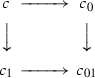(2.2)

in $$\mathcal {C}$$ defines an object $$\widetilde{c} \in \mathcal {C}^\mathsf{V}$$, $$\widetilde{c}(i)=c_i$$, $$i=0,1,01$$, and a map $$t:\tau (c) \rightarrow \widetilde{c}$$. Then the square is homotopy-cartesian if $$\langle \mathcal {C}^\mathsf{V},W^I \rangle$$ is localizable, and the map h(t) identifies h(c) with $$\mathsf{holim}_Ih(\widetilde{c})$$. A commutative square in $${\text {Ho}}(\mathcal {C},W)$$ is homotopy-cartesian if it is isomorphic to the image of a homotopy-cartesian square (2.2) in $$\mathcal {C}$$. Dually, a square in $$\mathcal {C}$$ resp. $${\text {Ho}}(\mathcal {C},W)$$ is homotopy-cocartesian if it is homotopy-cartesian in $$\mathcal {C}^o$$ resp. $${\text {Ho}}(\mathcal {C}^o,W^o)$$.

### Model structures

Our notion of a model category is that of  (called “closed model category” there). To fix terminology, here is the definition.

### Definition 2.6

A model structure on a saturated relative category $$\langle \mathcal {C},W \rangle$$ consists of two additional classes of maps C, F such that the following conditions are satisfied:

1. (i)

$$\mathcal {C}$$ has finite limits and colimits,

2. (ii)

C and F are closed under retracts and compositions,

3. (iii)

C has a left-lifting property with respect to $$F \cap W$$, and $$C \cap W$$ has a left-lifting property with respect to F, in the sense of , and

4. (iv)

every morphism g in $$\mathcal {C}$$ decomposes as $$g = f_1 \circ c_1 = f_1 \circ c_2$$, $$f_1 \in F$$, $$f_2 \in F \cap W$$, $$c_1 \in C \cap W$$, $$c_2 \in C$$.

A model category is a saturated relative category equipped with a model structure.

We will assume known standard terminology from  (in particular, maps in C, F, W are “cofibrations”, “fibrations”, “weak equivalences”, and an object $$X \in \mathcal {C}$$ is fibrant resp. cofibrant iff $$X \rightarrow 1$$ is a fibration resp. $$0 \rightarrow C$$ is a cofibration). We will also assume known the main results of  (for a very good exposition, see also ). In particular, one knows that a relative category $$\langle \mathcal {C},W \rangle$$ that admits a model structure is localizable, and any map in $${\text {Ho}}(\mathcal {C},W)$$ can be represented by an expression (2.1) of length at most 3. Every object in $${\text {Ho}}(\mathcal {C},W)$$ can be represented by an object $$X \in \mathcal {C}$$ that is fibrant and cofibrant, and for two such objects $$X,X' \in \mathcal {C}$$, any map $$f:h(X') \rightarrow h(X)$$ can be represented by a single map $$f:X' \rightarrow X$$. Two maps $$f_1,f_2:X' \rightarrow X$$ represent the same map in $${\text {Ho}}(\mathcal {C},W)$$ if and only if, for some fixed decomposition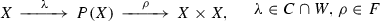(2.3)

of the diagonal embedding $$X \rightarrow X \times X$$, there exists a map $$f:X' \rightarrow P(X)$$ such that $$f_1 = \pi _1 \circ f$$ and $$f_2 = \pi _2 \circ f$$, where $$\pi _1,\pi _2:P(X) \rightarrow X$$ are the compositions of $$\rho$$ with the projections $$X \times X \rightarrow X$$ onto the first resp. the second factor. Both $$\pi _1$$ and $$\pi _2$$ are in $$F \cap W$$.

### Definition 2.7

Assume given a relative category $$\langle C,W \rangle$$ equipped with a model structure $$\langle C,F \rangle$$, and a small category I. A model structure $$\langle \widetilde{C},\widetilde{F} \rangle$$ on $$\langle \mathcal {C}^I,W^I \rangle$$ is injective if $$\widetilde{C} = C^I$$ and projective if $$\widetilde{F}=F^I$$.

We assume known that a model structure on a relative category $$\langle \mathcal {C},W \rangle$$ is completely defined by either of the two classes C, F (F consists of morphisms that have the right lifting property with respect to $$C \cap W$$, and C consists of morphisms that have the left lifting property with respect to $$F \cap W$$). Therefore the injective and projective model structures on $$\mathcal {C}^I$$ are unique, if they exist. In general, they do not. One situation where they do exist is when I is the category $$\mathsf{V}$$ used in the definition of homotopy cartesian and cocartesian squares. For any relative category $$\langle \mathcal {C},W \rangle$$ equipped with a model structure, the relative category $$\langle \mathcal {C}^\mathsf{V},W^\mathsf{V}\rangle$$ has the injective model structure, and $$\mathsf{holim}_\mathsf{V}E$$ exists for any $$E \in {\text {Ho}}(\mathcal {C}^\mathsf{V},W^\mathsf{V})$$. Dually, $$\langle \mathcal {C}^o,W^o \rangle$$ has a model structure (with $$F^o$$ as cofibrations and $$C^o$$ as fibrations), and $$\mathsf{hocolim}_{\mathsf{V}^o} E$$ exists for any $$E:\mathsf{V}^o \rightarrow \mathcal {C}$$. To represent homotopy cartesian and cocartesian squares in $$\mathcal {C}$$, it is convenient to introduce the following.

### Definition 2.8

A cofiber square in a model category $$\mathcal {C}$$ is a cocartesian square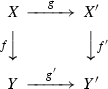(2.4)

in $$\mathcal {C}$$ such that X is cofibrant and $$f,g \in C$$. A fiber square in $$\mathcal {C}$$ is a cartesian square (2.4) such that $$Y'$$ is fibrant and $$f',g' \in F$$ (or equivalently, the square is a cofiber square in $$\mathcal {C}^o$$).

Then every homotopy cocartesian square in $$\mathcal {C}$$ is weakly equivalent to a cofiber square, and conversely, every cofiber square is homotopy cocartesian. In fact, a cocartesian square (2.4) is homotopy cocartesian as soon as X is cofibrant, and either f or g is in C (for a proof, see e.g.  or Sect. 7.2). For fiber squares, the situation is dual.

For any integer $$n \ge 0$$, denote by [n] the totally ordered set $$\{0,\dots ,n\}$$ considered as a small category in the usual way. Then for any model structure on a relative category $$\langle \mathcal {C},W \rangle$$, the category $$\langle \mathcal {C}^{[n]},W^{[n]} \rangle$$ also has the injective and projective model structures. We will need the following simple lemma.

### Lemma 2.9

For any relative category $$\langle \mathcal {C},W \rangle$$ equipped with a model structure, and any integer $$n \ge 1$$, the tautological functor

\begin{aligned} \varphi :{\text {Ho}}(\mathcal {C}^{[n]},W^{[n]}) \rightarrow {\text {Ho}}(\mathcal {C},W)^{[n]} \end{aligned}

is essentially surjective and full.

### Proof

Essential surjectivity is obvious (represent the values of a functor $$[n] \rightarrow \mathcal {C}$$ by fibrant cofibrant objects, and lift the maps between adjacent values in any way). To prove that $$\varphi$$ is full, assume given diagrams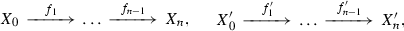representing objects $$X_\cdot ,X'_\cdot \in \mathcal {C}^{[n]}$$, and a map $$g:\varphi (h(X'_\cdot )) \rightarrow \varphi (h(X_\cdot ))$$ given by a collection of maps $$g_i:h(X_i') \rightarrow h(X_i)$$ such that $$h(f_i) \circ g_i = g_{i+1} \circ h(f'_i)$$, $$i = 1,\dots ,n-1$$. To prove the claim, it suffices to lift $$g_\cdot$$ to maps $$\widetilde{g}_\cdot :X_\cdot ' \rightarrow X_\cdot$$ such that $$f_\cdot \circ g_\cdot = g_{\cdot +1} \circ f'_\cdot$$.

We may assume that $$X_\cdot$$ and $$X'_\cdot$$ are fibrant and cofibrant for the projective model structure on $$\mathcal {C}^{[n]}$$, so that $$f_\cdot ,f'_\cdot \in C$$. Moreover, we may assume by induction that we already have the maps $$\widetilde{g}_i$$ for $$i = 0,\dots ,n-1$$.

Choose a decomposition (2.3) for the object $$X_n \in \mathcal {C}$$, and take an arbitrary map $$g:X_n' \rightarrow X_n$$ such that $$h(g)=g_n$$. Then $$h(g \circ f_{n-1}') = h(f_{n-1} \circ \widetilde{g}_{n-1})$$, so that there exists a map $$\widetilde{g}:X'_{n-1} \rightarrow P(X_n)$$ such that $$g \circ f_{n-1}' = \pi _1 \circ \widetilde{g}$$ and $$f_{n-1} \circ \widetilde{g}_{n-1} = \pi _2 \circ \widetilde{g}$$. But $$\pi _1 \in F \cap W$$, so by the lifting property, there exists a map $$q:X'_n \rightarrow P(X_n)$$ such that $$\widetilde{g} = q \circ f'_{n-1}$$ and $$g = \pi _1 \circ q$$. Take $$\widetilde{g}_n = \pi _2 \circ q$$, and note that $$h(\widetilde{g}_n) = h(g) = g_n$$, and $$\widetilde{g}_n \circ f'_{n-1} = \pi _2 \circ q = f_{n-1} \circ \widetilde{g}_{n-1}$$. $$\square$$

Finally, we make one general remark. If one takes a relative category $$\langle \mathcal {C},W \rangle$$ as a primary object of study, then a model structure in the sense of Definition 2.6 is a choice. Making this choice ensures that $$\langle \mathcal {C},W \rangle$$ is localizable and $${\text {Ho}}(\mathcal {C},W)$$ is well-behaved, but $${\text {Ho}}(\mathcal {C},W)$$ itself does not depend on the choice made. Unfortunately, the choice is not always possible—for example, many relative categories needed in applications do not satisfy Definition 2.6 (i). To alleviate the problem, it is convenient to introduce the following.

### Definition 2.10

A model embedding of a relative category $$\langle \mathcal {C},W \rangle$$ is a fully faithful functor $$\langle \mathcal {C},W \rangle \rightarrow \langle \mathcal {C}',W' \rangle$$ to a relative category $$\langle \mathcal {C}',W' \rangle$$ equipped with a model structure such that for any weak equivalence $$w:X \rightarrow X'$$, $$w \in W'$$, we have $$X \in \mathcal {C}\subset \mathcal {C}'$$ if and only if $$X' \in \mathcal {C}\subset \mathcal {C}'$$.

### Lemma 2.11

If a model category $$\langle \mathcal {C},W \rangle$$ admits a model embedding $$\varepsilon :\langle \mathcal {C},W \rangle \rightarrow \langle \mathcal {C}',W \rangle$$, then $$\langle \mathcal {C},W \rangle$$ is localizable, and $${\text {Ho}}(\varepsilon ):{\text {Ho}}(\mathcal {C},W) \rightarrow {\text {Ho}}(\mathcal {C}',W')$$ is fully faithful.

### Proof

Assume given two objects $$X_0,X_1 \in \mathcal {C}\subset \mathcal {C}'$$, and chose a cofibrant replacement $$w_0:X_0' \rightarrow X_0$$ and a fibrant replacement $$w_1:X_1 \rightarrow X_1'$$. Then by the standard argument of , any diagram (2.1) in $$\mathcal {C}'$$ representing a map from $$X_0$$ to $$X_1$$ in $${\text {Ho}}(\mathcal {C}',W')$$ can be reduced to $$w_1^{-1} \circ f \circ w_0^{-1}$$ for some map $$f:X_0' \rightarrow X_1'$$. But since $$\varepsilon$$ is a model embedding, both $$X_0'$$ and $$X_1'$$ lie in $$\mathcal {C}\subset \mathcal {C}'$$, and moreover, the same reduction works entirely within $$\mathcal {C}$$. This insures that $${\text {Ho}}(\mathcal {C},W)(X_0,X_1)$$ is a quotient of the set $$\mathcal {C}(X_0',X_1')$$, thus itself a set, so that $$\langle \mathcal {C},W \rangle$$ is localizable. Moreover, $${\text {Ho}}(\varepsilon ):{\text {Ho}}(\mathcal {C},W) \rightarrow {\text {Ho}}(\mathcal {C}',W')$$ is full. To finish the proof, it remains to show that if for some maps $$f,f':X_0' \rightarrow X_1'$$ we have $$w_1^{-1} \circ f \circ w_0^{-1} = w_1^{-1} \circ f \circ w_0^{-1}$$ in $${\text {Ho}}(\mathcal {C}',W')$$, then the same holds on $${\text {Ho}}(\mathcal {C},W)$$. But by Quillen , the expressions are equal in $${\text {Ho}}(\mathcal {C}',W')$$ if and only if $$f = h \circ g$$, $$f' = h \circ g'$$ for some map $$\widetilde{f}:Y \rightarrow X_1$$, where $$\widetilde{f}$$ is obtained by fixing a factorization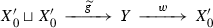of the codiagonal map $$X_0' \sqcup X_0' \rightarrow X_0'$$, with $$\widetilde{g} \in C$$, $$w \in F \cap W$$, and g, $$g'$$ are the compositions of the map $$\widetilde{g}$$ with the two embeddings $$X_0' \rightarrow X_0' \sqcup X_0'$$. Since in this case, Y is also in $$\mathcal {C}\subset \mathcal {C}'$$, we indeed have $$w_1^{-1} \circ f \circ w_0^{-1} = w_1^{-1} \circ f \circ w_0^{-1}$$ in $${\text {Ho}}(\mathcal {C},W)$$. $$\square$$

By virtue of Lemma 2.11, while $$\langle \mathcal {C},W \rangle$$ itself does not necessarily have a model structure, it can still be studied by model category techniques. From the general point of view, this is only logical—if we need to choose a model structure anyway, why not choose also an ambient category that can carry it with some comfort.

### Stability

Recall that a category $$\mathcal {C}$$ is called pointed if it has an initial object 0 and a terminal object 1, and the unique map $$0 \rightarrow 1$$ is an isomorphism. Given a pointed category $$\mathcal {C}$$, we denote the initial terminal object by 0, so that for any $$X \in \mathcal {C}$$ we have a unique map $$0 \rightarrow X$$ and a unique map $$X \rightarrow 0$$ (both maps are also denoted by 0). If a pointed category $$\mathcal {C}$$ has finite products and coproducts, then for any two objects $$X,Y \in \mathcal {C}$$, we have a natural map

\begin{aligned} X \sqcup Y \rightarrow X \times Y, \end{aligned}
(2.5)

the coproduct of the map $$\mathsf{id}\times 0$$ and the map $$0 \times \mathsf{id}$$.

If a localizable relative category $$\langle \mathcal {C},W \rangle$$ is pointed, then $${\text {Ho}}(\mathcal {C},W)$$ is also pointed, with the same object 0. We will say that $$X \in \mathcal {C}$$ is acyclic if $$h(X) \cong 0$$ (equivalently, $$X \rightarrow 0$$ is in W, equivalently, $$0 \rightarrow X$$ is in W).

Assume given a pointed relative category $$\langle \mathcal {C},W \rangle$$ equipped with a model structure. Then by definition, $$\mathcal {C}$$ has finite sums and products, and these descend to $${\text {Ho}}(\mathcal {C},W)$$. Moreover, consider the category $$\mathcal {C}^{}$$ (that is, the category of arrows $$f:X \rightarrow Y$$ in $$\mathcal {C}$$). We have two functors $$\widetilde{S},\widetilde{T}:\mathcal {C}\rightarrow \mathcal {C}^{}$$ sending $$X \in \mathcal {C}$$ to $$X \rightarrow 0$$ resp. $$0 \rightarrow X$$, and these descend to functors

\begin{aligned} S,T:{\text {Ho}}(\mathcal {C},W) \rightarrow {\text {Ho}}(\mathcal {C}^{},W^{}). \end{aligned}

The functor $$\widetilde{S}$$ is left-Quillen with respect to the injective model structure on $$\mathcal {C}^{}$$, and the functor $$\widetilde{T}$$ is right-Quillen with respect to the projective model structure. Therefore we have natural functors

\begin{aligned} S^\dagger ,T_\dagger :{\text {Ho}}(\mathcal {C}^{},W^{}) \rightarrow {\text {Ho}}(\mathcal {C},W), \end{aligned}
(2.6)

$$S^\dagger$$ adjoint to S on the right, and $$T_\dagger$$ adjoint to T on the left. Any commutative square (2.4) in $$\mathcal {C}$$ with acyclic $$Y'$$ induces a natural map $$T_\dagger (h(f)) \rightarrow h(X')$$, and this map is an isomorphism if and only if (2.4) is homotopy cocartesian. This holds for example if the square is cocartesian and $$f \in C$$. Analogously, (2.4) with acyclic Y induces a map $$h(X) \rightarrow S^\dagger (h(f))$$, the map is an isomorphism if and only if (2.4) is homotopy cartesian, and this holds in particular if the square is cartesian and $$f' \in F$$. We also note that a square (2.4) with acyclic X induces a map $$h(X') \sqcup h(Y) \rightarrow Y'$$, this map is an isomorphism if and only if (2.4) is homotopy cocartesian, and dually for a square with acyclic $$Y'$$.

Composing the functors S, T with the functors $$T_\dagger$$, $$S^\dagger$$, we obtain a pair of functors

\begin{aligned} \Sigma = T_\dagger \circ S,\Omega = S^\dagger \circ T:{\text {Ho}}(\mathcal {C}) \rightarrow {\text {Ho}}(\mathcal {C}), \end{aligned}
(2.7)

with $$\Sigma$$ adjoint to $$\Omega$$ on the left.

### Definition 2.12

A stable model pair is a pair of a pointed relative category $$\langle \mathcal {C},W \rangle$$ and a pointed model embedding $$\langle \mathcal {C},W \rangle \rightarrow \langle \mathcal {C}',W' \rangle$$ such that

1. (i)

a square (2.4) with $$X,Y \in \mathcal {C}$$ is homotopy cartesian if and only if it is homotopy cocartesian, and

2. (ii)

in this case, $$X' \in \mathcal {C}$$ if and only if $$Y' \in \mathcal {C}$$.

### Example 2.13

Assume given an abelian category $$\mathcal {C}$$, let $$C_\cdot (\mathcal {C})$$ be the category of chain complexes in $$\mathcal {C}$$, and let W be the class of quasiisomorphisms. Then if $$\mathcal {C}$$ has enough injectives, $$C_\cdot (\mathcal {C})$$ has a model structure such that C consists of termwise-injective maps, called the injective model structure, and if $$\mathcal {C}$$ has enough projectives, it has the projective model structure with F formed by termwise-surjective maps. Both are stable. If we have an abelian subcategory $$\mathcal {C}_0 \subset \mathcal {C}$$—for example, coherent sheaves on a Noetherian scheme inside of quasicoherent schemes—and $$C_\cdot (\mathcal {C})$$ has either the injective or the projective model structure, then $$\langle C_\cdot (\mathcal {C}_0),C_\cdot (\mathcal {C}) \rangle$$ is a stable model pair.

Without the model embedding part, Definition 2.12 gives the very standard notion of a stable model category whose application to triangulated categories goes back at least to . As it happens, most of the theory carries over to stable model pairs. We prove several simple results right away.

### Lemma 2.14

For any stable model pair $$\langle \mathcal {C},W \rangle \subset \langle \mathcal {C}',W' \rangle$$, the functors $$\Sigma$$, $$\Omega$$ of (2.7) send $${\text {Ho}}(\mathcal {C}) \subset {\text {Ho}}(\mathcal {C}')$$ to itself and induce adjoint autoequivalences of $${\text {Ho}}(\mathcal {C})$$.

### Proof

Any object in $${\text {Ho}}(\mathcal {C})$$ can be represented by a cofibrant object $$X \in \mathcal {C}\subset \mathcal {C}'$$, and we can further choose a cofibration $$f:X \rightarrow Y$$ with acyclic Y. Extend f to a cofiber square (2.4) in the sense of Definition 2.8, say with $$X = Y$$. Then f represents S(h(X)) in $${\text {Ho}}(\mathcal {C}^{},W^{})$$, and being cocartesian, (2.4) induces an isomorphism $$\Sigma (h(X)) \cong h(Y')$$. Since $$X=Y$$ is an acyclic object, and $$\mathcal {C}\subset \mathcal {C}'$$ is a pointed model embedding, $$X=Y$$ lies in $$\mathcal {C}$$. Then by Definition 2.12 (ii), $$Y' \in \mathcal {C}$$, and by Definition 2.12 (i), (2.4) is homotopy cartesian, thus induces an isomorphism $$h(X) \cong \Omega (h(Y'))$$. Therefore $$\Sigma ({\text {Ho}}(C,W)) \subset {\text {Ho}}(\mathcal {C},W)$$, and the adjunction map $$h(X) \rightarrow \Omega (\Sigma (h(X)))$$ is an isomorphism for any $$X \in \mathcal {C}$$. By the dual argument, $$\Omega ({\text {Ho}}(\mathcal {C},W)) \subset {\text {Ho}}(\mathcal {C},W)$$, and the adjunction map $$\Sigma (\Omega (h(X))) \rightarrow h(X)$$ is also an isomorphism for any $$X \in \mathcal {C}$$. $$\square$$

### Lemma 2.15

For any stable model pair $$\langle \mathcal {C},W \rangle \subset \langle \mathcal {C}',W' \rangle$$, the category $${\text {Ho}}(\mathcal {C},W)$$ has finite products and coproducts, and the map (2.5) is an isomorphism for any $$X,Y \in {\text {Ho}}(\mathcal {C},W)$$.

### Proof

Since $$\langle \mathcal {C}',W' \rangle$$ has a model structure, $${\text {Ho}}(\mathcal {C}',W')$$ has products and coproducts, and $${\text {Ho}}(\mathcal {C},W) \subset {\text {Ho}}(\mathcal {C}',W')$$ is closed under products and coproducts by Definition 2.12 (ii). For the second claim, take two objects in $${\text {Ho}}(\mathcal {C},W)$$, represent them by fibrant objects $$X,Y \in \mathcal {C}$$, and construct a commutative diagram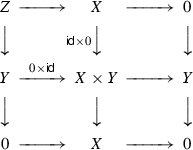with homotopy cartesian top-left square. The other three squares are cartesian, and since X, Y are fibrant, they are homotopy cartesian. Therefore the outer square is also homotopy cartesian, so that Z is acyclic, and if we replace it with 0, the top-left square is still homotopy cartesian. By Definition 2.12 (i), it is then also homotopy cocartesian, and this exactly means that (2.5) is an isomorphism. $$\square$$

By Lemma 2.15, for any two objects $$X,Y \in {\text {Ho}}(\mathcal {C})$$, we can from now on denote $$X \sqcup Y \cong X \times Y$$ by $$X \oplus Y$$. Moreover, the set $${\text {Ho}}(\mathcal {C})(X,Y)$$ of maps between X and Y has a natural structure of a commutative monoid, with $$X \rightarrow 0 \rightarrow Y$$ being the unit, and the sum $$f+f'$$ of two maps $$f,f':X \rightarrow Y$$ given by the composition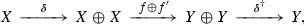where $$\delta$$ is the diagonal map, and $$\delta ^\dagger$$ is the codiagonal map.

For our final result, consider a cofibrant object $$X \in \mathcal {C}$$, and form a cofiber square (2.4) with acyclic Y and $$X'$$. Then it defines an isomorphism $$l:h(Y') \cong \Sigma (h(X))$$. On the other hand, we can flip the square and consider it as a map from its top row to its bottom row. This also gives a canonical isomorphism $$r:h(Y') \cong \Sigma (h(X))$$.

### Lemma 2.16

For any stable model pair $$\langle \mathcal {C},W \rangle \subset \langle \mathcal {C}',W' \rangle$$ and any cofiber square (2.4) in $$\mathcal {C}'$$ with $$X \in \mathcal {C}$$ and acyclic Y, $$X'$$, the isomorphisms $$l,r:h(Y') \cong \Sigma (h(X))$$ satisfy $$l+r=0$$.

### Proof

Since all cofiber squares (2.4) with acyclic Y, $$X'$$ are weakly equivalent, the claim does not depend on the choice of the square. Choose one with $$X'=Y$$ and the same cofibration $$f:X \rightarrow Y$$ on the left and on top. Define $$\Sigma (X)$$ by the cocartesian square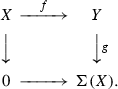Since f is a cofibration, $$h(\Sigma (X)) \cong \Sigma (h(X))$$, and the map $$l + r = \delta ^\dagger \circ (l \oplus r)$$ can be obtained by considering the commutative diagram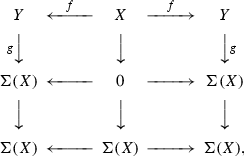(2.8)

treating its rows as objects in $$\mathcal {C}^\mathsf{V}$$, and taking $$\mathsf{hocolim}_\mathsf{V}$$. It remains to observe that the resulting map from the top row to the bottom row of (2.8) factors through the object $$Y \leftarrow Y \rightarrow Y$$ in $$\mathcal {C}^\mathsf{V}$$, and this object is acyclic. $$\square$$

### Corollary 2.17

The category $${\text {Ho}}(\mathcal {C})$$ is additive.

### Proof

By Lemma 2.15, $${\text {Ho}}(\mathcal {C})$$ has finite sums and products that coincide, and spaces of maps in $${\text {Ho}}(\mathcal {C})$$ are commutative monoids. It remains to check that they are abelian groups. Indeed, by Lemma 2.16, we have an automorphism $$\sigma :\Sigma \rightarrow \Sigma$$ of the functor $$\Sigma$$ such that $$\mathsf{Id}+ \sigma = 0$$, so that any $$f:X \rightarrow Y$$ has an inverse element $$-f = \Omega (\sigma \circ \Sigma (f))$$. $$\square$$

### Triangulated structures

The main property of stable model pairs is that they produce triangulated categories. To state this, let us recall the precise definition.

### Definition 2.18

A triangulated category is an additive category T equipped with an autoequivalence $$X \mapsto X$$ and a class of distinguished triangles of the form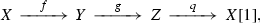(2.9)

satisfying the following axioms:

1. (TR1)

For any $$X \in T$$, the triangle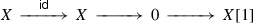is distinguished. A triangle (2.9) isomorphic to a distinguished one is itself distinguished, and any map $$f:X \rightarrow Y$$ fits into a distinguished triangle (2.9).

2. (TR2)

For any distinguished triangle (2.9), the triangle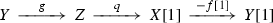is distinguished.

3. (TR3)

For any distinguished triangle (2.9), another distinguished triangle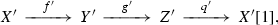and two maps $$x:X \rightarrow X'$$, $$y:Y \rightarrow Y'$$ such that $$y \circ f = f' \circ x$$, there exists a map $$z:Z \rightarrow Z'$$ such that $$z \circ g = g' \circ y$$ and $$x \circ q = q' \circ z$$.

4. (TR4)

For any three objects $$X,Y,Z \in T$$ and two maps $$f:X \rightarrow Y$$, $$g:Y \rightarrow Z$$, there exists a commutative diagram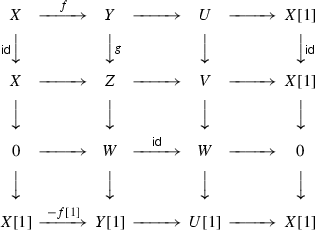(2.10)

with distinguished rows and columns.

Formally, Definition 2.18 (TR4) is weaker than the octahedron axiom of . However, as proved for instance in [19, Chapter IV, §1.4], the axioms (TR1)-(TR3) imply that a distinguished triangle (2.9) is defined by $$f:X \rightarrow Y$$ up to a (non-unique) isomorphism; this together with (TR4) is equivalent to the octahedron axiom in its usual form.

### Remark 2.19

From time to time, people try to prove that (TR4) itself follows from the other axioms, or alternatively, that it can be dispensed with altogether without any noticable ill effects. One of the reasons for this is that historically, the first definition of a triangulated category in the literature appeared in , and the axiom (TR4) was not included. Let us mention that nevertheless, at least from the perspective of , this is a very strange way to look at things. Indeed, the whole point of introducing Definition 2.18 in  was that it has a non-trivial theorem attached to it—namely, the localization theorem that constructs the quotient $${\mathcal {D}}/{\mathcal {D}}_0$$ of a triangulated category $${\mathcal {D}}$$ by a triangulated subcategory $${\mathcal {D}}_0$$ (we recall the precise formulation in Sect. 3.4). For this purpose, (TR4) is the most important axiom: it insures that the class of morphisms in $${\mathcal {D}}$$ whose cone lies in $${\mathcal {D}}_0$$ is saturated in the sense of Definition 2.2, and gives one control over the localization process.

Assume now given a stable model pair $$\langle \mathcal {C},W \rangle \subset \langle \mathcal {C}',W' \rangle$$ in the sense of Definition 2.12. By Lemma 2.14, setting $$X = \Sigma (X)$$ defines an autoequivalence of the category $${\text {Ho}}(\mathcal {C})$$. For any cofibration $$f:X \rightarrow Y$$ of cofibrant objects in $$\mathcal {C}$$, choose cofibrations $$X \rightarrow A$$, $$Y \rightarrow B$$ with acyclic A, B, and form the cofiber squares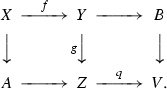(2.11)

Then the outer rectangle is also a cofiber square, so that we obtain an identification $$h(V) \cong h(X)$$ and a triangle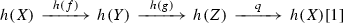(2.12)

in $${\text {Ho}}(\mathcal {C})$$. Say that a triangle (2.9) in $${\text {Ho}}(\mathcal {C})$$ is distinguished if it is isomorphic to the triangle (2.12) induced by a diagram (2.11).

### Proposition 2.20

The category $${\text {Ho}}(\mathcal {C})$$ with the shift $$X \mapsto X$$ and the class of distinguished triangles defined above is a triangulated category in the sense of Definition 2.18.

At least when $$\mathcal {C}=\mathcal {C}'$$, this fact is extremely well-known since at least , but short written proofs are not easy to come by. In  much more is proved, but extracting exactly the claim of Proposition 2.20 is a rather non-trivial exercise (one should also keep in mind the fact that in , triangulated categories are called “classical triangulated categories”, and a triangulated category in the sense [26, Definition 7.1.1] has a form of enhancement already hardcoded into it). More recently, a proof in the $$\infty$$-categorical setting appears in , and a complete and detailed proof in the setting of derivators is worked out in . However, just as [24, 26] proves much more than what we claim here, so the paper is rather long, and on the other hand, it still does not cover the case of model pairs. So we think that giving a short and self-contained proof is useful. We emphasize that the argument is still the same, and claim no conceptual novelty whatsoever.

### Proof of Proposition 2.20

The category $${\text {Ho}}(\mathcal {C},W)$$ is additive by Corollary 2.17. All the claims of (TR1) except for the last one hold by definition, and the last claim follows from the first claim of Lemma 2.9 for $$n=2$$. The second claim of Lemma 2.9 for $$n=2$$ gives (TR3). For (TR2), consider a diagram (2.11), choose a cofibration $$Z \rightarrow C$$ with acyclic C, and extend (2.11) to a diagram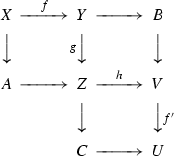with three cofiber squares. Then the right-hand side part of this diagram is a flipped diagram of the form (2.11). This gives identifications $$h(V) \cong h(X)$$, $$h(U) \cong h(Y)$$, and Lemma 2.16 then shows that under these identifications, we have $$f' + f = 0$$. Finally, for (TR4), note that any object in $$\mathcal {C}^{}$$ automatically generates a diagram (2.10); the claim then immediately follows from the first claim of Lemma 2.9 for $$n=3$$. $$\square$$

### Remark 2.21

Note that both stable model pairs and triangulated structures are self-dual notions, so that Proposition 2.20 actually provides two triangulated structures on $${\text {Ho}}(\mathcal {C},W)^o \cong {\text {Ho}}(\mathcal {C}^o,W^o)$$. The triangles in the second structure are generated by diagrams (2.11) with fiber squares instead of cofiber squares. However, Definition 2.12 (i) immediately shows that the two structures coincide.

### Lemma 2.22

Assume given a stable model pair $$\langle \mathcal {C},\mathcal {C}' \rangle$$ and a full triangulated subcategory $${\mathcal {D}}_0 \subset {\mathcal {D}}= {\text {Ho}}(\mathcal {C})$$, and let $$\mathcal {C}_0 \subset \mathcal {C}$$ be the full subcategory spanned by objects X with $$h(X) \in {\mathcal {D}}_0$$. Then $$\langle \mathcal {C}_0,\mathcal {C}' \rangle$$ is a stable model pair.

### Proof

By definition, $$\mathcal {C}_0 \subset \mathcal {C}'$$ is a model embedding, and Definition 2.12 (i) follows from the corresponding property of $$\mathcal {C}\subset \mathcal {C}'$$. For Definition 2.12 (ii), note that the fourth term in a homotopy cartesian or cocartesian square (2.4) can be expressed as a cone of a map between sums of shifts of the other three terms, and $${\mathcal {D}}\subset {\text {Ho}}(\mathcal {C})$$ is by assumption stable under cones, shifts and finite sums. $$\square$$

## Elementary gluing

### Functors

The standard class of functors between model categories that induce natural derived functors on the homotopy level are the left and right-Quillen functors. However, for some applications this notion is too strong. Therefore let us introduce the following.

### Definition 3.1

A functor $$\Phi :\langle \mathcal {C}_0,W_0 \rangle \rightarrow \langle \mathcal {C}_1,W_1 \rangle$$ between two relative categories with model structures $$C_0,F_0$$ and $$C_1,F_1$$ is left-derivable if it sends morphisms in $$C_0$$ to morphisms in $$C_1$$ and morphisms in $$C_0 \cap W_0$$ to morphisms in $$C_1 \cap W_1$$. A functor $$\Phi$$ is right-derivable if $$\Phi ^o$$ is left-derivable.

The difference between left-derivable functors of Definition 3.1 and left-Quillen functors is exactness: we do not require our functors to preserve finite colimits. Still, as in , any left-derivable functor $$\Phi :\mathcal {C}_0 \rightarrow \mathcal {C}_1$$ induces a natural left-derived functor $$L^\cdot \Phi :{\text {Ho}}(\mathcal {C}_0,W_0) \rightarrow {\text {Ho}}(\mathcal {C}_1,W_1)$$ obtained by restricting $$\Phi$$ to the full subcategory spanned by cofibrant objects in $$\mathcal {C}_0$$. Analogously, a right-derivable functor $$\Phi$$ induces a right-derived functor $$R^\cdot \Phi :{\text {Ho}}(\mathcal {C}_0,W_0) \rightarrow {\text {Ho}}(\mathcal {C}_1,W_1)$$. In these terms, the Quillen Adjunction Theorem can be restated as follows: if $$\Phi ^\dagger$$ is right-adjoint to $$\Phi$$, and $$\Phi$$ is left-derivable, then $$\Phi ^\dagger$$ is right-derivable, and $$R^\cdot \Phi ^\dagger$$ is right-adjoint to $$L^\cdot \Phi$$. Another trivial but useful observation is the following: if a functor $$\Phi :\mathcal {C}_0 \rightarrow \mathcal {C}_1$$ is both left and right-derivable, then it sends W to W, thus induces a functor $${\text {Ho}}(\Phi ):{\text {Ho}}(\mathcal {C}_0) \rightarrow {\text {Ho}}(\mathcal {C}_1)$$, and we have $$L^\cdot \Phi \cong {\text {Ho}}(\Phi ) \cong R^\cdot \Phi$$.

### Definition 3.2

Assume given stable model pairs $$\langle \mathcal {C}_0',\mathcal {C}_0 \rangle$$, $$\langle \mathcal {C}_1',\mathcal {C}_1 \rangle$$. A left resp. right-derivable functor $$\Phi :\mathcal {C}_0 \rightarrow \mathcal {C}_1$$ is stable if it is pointed, and $$L^\cdot \Phi$$ resp. $$R^\cdot \Phi$$ sends $${\text {Ho}}(\mathcal {C}_0') \subset {\text {Ho}}(\mathcal {C}_0)$$ into $${\text {Ho}}(\mathcal {C}_1') \subset {\text {Ho}}(\mathcal {C}_1)$$ and homotopy cartesian squares of Definition 2.12 (i) in $${\text {Ho}}(\mathcal {C}_0')$$ to homotopy cartesian squares in $${\text {Ho}}(\mathcal {C}_1')$$.

### Remark 3.3

By Definition 2.12 (i), replacing homotopy cartesian squares in Definition 3.2 with homotopy cocartesian squares gives the same notion.

### Remark 3.4

In the assumptions of Definition 3.2, a right-Quillen functor $$\Phi :\mathcal {C}_0 \rightarrow \mathcal {C}_1$$ is obviously stable as soon as $$R^\cdot \Phi$$ sends $${\text {Ho}}(\mathcal {C}_0')$$ into $${\text {Ho}}(\mathcal {C}_1')$$.

### Example 3.5

Assume given a left-exact functor $$\Phi :\mathcal {C}\rightarrow \mathcal {E}$$ between Grothendieck abelian categories. Then the induced functor $$\Phi _\cdot :C_\cdot (\mathcal {C}) \rightarrow C_\cdot (\mathcal {E})$$ is right-Quillen, hence also right-derivable with respect to the injective model structures, and $$R^\cdot \Phi _\cdot$$ is the usual derived functor of the functor $$\Phi$$.

However, it is certainly not true that all right-derivable functors between categories of complexes are of this type. For example, assume given algebraic varieties X, Y and a complex $$K \in {\mathcal {D}}^+_{coh}(X \times Y)$$ of sheaves on $$X \times Y$$ with coherent cohomology. Then we have the Fourier–Mukai functor $$\Phi :{\mathcal {D}}(X) \rightarrow {\mathcal {D}}(Y)$$ between the derived categories of quasicoherent sheaves on X and Y given by

\begin{aligned} \Phi (\mathcal {F}) = R^\cdot \pi _{2*}\left( L^\cdot \pi _1^*\mathcal {F}\overset{\mathsf{\scriptscriptstyle L}}{\otimes }K\right) , \end{aligned}

where $$\pi _1:X \times Y \rightarrow X$$, $$\pi _2:X \times Y \rightarrow Y$$ are the projections. In general, $$\Phi$$ is not the derived functor of any functor between the abelian categories. However, to realize it as $$R^\cdot \Phi _\cdot$$ for some right-Quillen functor $$\Phi _\cdot :C_\cdot (X) \rightarrow C_\cdot (Y)$$, it suffices to equip both categories with injective model structures, and represent K by a complex of coherent sheaves flat over X.

### Example 3.6

To see an example of a derivable functor that is not Quillen, consider the category $$C_\cdot (k)$$ of complexes of vector spaces over a field k. Since k is a field, all the natural model structures on $$C_\cdot (k)$$ coincide. Following , say that a sequence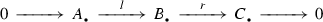in $$C_\cdot (k)$$ is quasiexact if $$r \circ l = 0$$, l is injective, r is surjective, and $$r/l$$ is an acyclic complex. Moreover, say that a pointed functor $$\Phi :C_\cdot (k) \rightarrow C_\cdot (k)$$ is admissible if it sends quasiexact sequences to quasiexact sequences (note that $$\Phi$$ is not required to be additive). Then an admissible functor $$\Phi$$ is both left and right-derivable with respect to the natural model structure in the sense of Definition 3.1. Moreover, $$\langle C_\cdot (k),C_\cdot (k) \rangle$$ is a stable model pair, and $$\langle \Phi ,\Phi \rangle$$ is stable in the sense of Definition 3.2.

### Lemma 3.7

For any stable left-derivable functor $$\Phi :\mathcal {C}_0 \rightarrow \mathcal {C}_1$$ between stable model pairs $$\langle \mathcal {C}_0',\mathcal {C}_0 \rangle$$ and $$\langle \mathcal {C}_1',\mathcal {C}_1 \rangle$$, the functor

\begin{aligned} L^\cdot \Phi :{\text {Ho}}\left( \mathcal {C}_0',W_0\right) \rightarrow {\text {Ho}}\left( \mathcal {C}_1',W_1\right) \end{aligned}

induced by the left-derived functor $$L^\cdot \Phi$$ is naturally triangulated with respect to the triangulated structures of Proposition 2.20. For a stable right-derivable functor $$\Phi$$, the same is true for the right-derived functor $$R^\cdot \Phi$$.

### Proof

By Remark 2.21, it suffices to consider the case of the left-derivable functor; for the right-derivable functor, everything then follows by passing to the opposite categories. By definition, every cofiber square (2.4) in $$\mathcal {C}_0'$$ induces a commutative square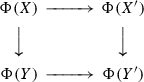in $$\mathcal {C}_1'$$, and since $$\Phi$$ is stable, the induced natural map $$\Phi (X') \sqcup _{\Phi (X')} \Phi (Y) \rightarrow \Phi (Y')$$ is a weak equivalence. Choosing a square with acylic $$X'$$, $$Y'$$, we then obtain an isomorphism

\begin{aligned} \Sigma (L^\cdot \Phi (h(X))) \cong L^\cdot \Phi (\Sigma (h(X))) \end{aligned}

that is obviously independent of the choices and functorial in X. This provides a functorial isomorphism $$L^\cdot \Phi (X) \cong L^\cdot \Phi (X)$$, $$X \in {\text {Ho}}(\mathcal {C}_0)$$. Moreover, by the same argument, if we apply $$\Phi$$ to a diagram (2.11) in $$\mathcal {C}_0$$ with cofiber squares, the result is weakly equivalent to a diagram (2.11) in $$\mathcal {C}_1$$ with cofiber squares, so that $$L^\cdot \Phi$$ is a triangulated functor. $$\square$$

### Simple gluing

Recall that for any functor $$\Phi :\mathcal {C}_0 \rightarrow \mathcal {C}_1$$ between two categories $$\mathcal {C}_0$$, $$\mathcal {C}_1$$, the right comma-category $$\mathsf{R}(\Phi )$$ is the category of triples $$\langle c_0,c_1,\alpha \rangle$$ of an object $$c_0 \in \mathcal {C}_0$$, an object $$c_1 \in \mathcal {C}_1$$, and a map $$\alpha :c_1 \rightarrow \Phi (c_0)$$. Morphisms from $$\langle c_0,c_1,\alpha \rangle$$ to $$\langle c_0',c_1',\alpha ' \rangle$$ are given by pairs $$\langle f_0,f_1 \rangle$$ of maps $$f_0:c_0 \rightarrow c_0'$$, $$f_1:c_1 \rightarrow c_1'$$ such that the square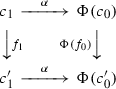(3.1)

is commutative. We have natural projections

\begin{aligned} \sigma :\mathsf{R}(\Phi ) \rightarrow \mathcal {C}_0, \qquad \tau :\mathsf{R}(\Phi ) \rightarrow \mathcal {C}_1 \end{aligned}
(3.2)

sending $$\langle c_0,c_1,\alpha \rangle$$ to $$c_0$$ resp. $$c_1$$, and the functor $$\sigma$$ has a right-adjoint $$\mathcal {C}_0 \rightarrow \mathsf{R}(\Phi )$$ sending $$c_0$$ to $$\langle c_0,\Phi (c_0),\mathsf{id}\rangle$$. The left comma-category $$\mathsf{L}(\Phi )$$ is given by $$\mathsf{L}(\Phi ) = \mathsf{R}(\Phi ^o)^o$$. Explicitly, its objects are triples $$\langle c_0,c_1,\alpha \rangle$$, $$c_\cdot \in \mathcal {C}_\cdot$$, $$\alpha :\Phi (c_0) \rightarrow c_1$$, we still have projections (3.2), and sending $$c_0$$ to $$\langle c_0,\Phi (c_0),\mathsf{id}\rangle$$ gives a left-adjoint to the projection $$\sigma$$.

### Proposition 3.8

1. (i)

Assume given a functor $$\Phi :\mathcal {C}_0 \rightarrow \mathcal {C}_1$$ between two model categories that is right-derivable in the sense of Definition 3.1. Then the comma-category $$\mathsf{R}(\Phi )$$ has a (unique) model structure such that $$\langle f_0,f_1 \rangle$$ lies in W resp. C if and only if so do $$f_0$$ and $$f_1$$. Moreover, if $$\langle f_0,f_1 \rangle$$ is in F with respect to this model structure, then so are $$f_0$$ and $$f_1$$.

2. (ii)

Assume given stable model pairs $$\langle \mathcal {C}_0',\mathcal {C}_0 \rangle$$, $$\langle \mathcal {C}_1',\mathcal {C}_1 \rangle$$, assume that $$\Phi$$ is stable in the sense of Definition 3.2, and let $$\mathsf{R}(\Phi )' \subset \mathsf{R}(\Phi )$$ the full subcategory spanned by triples $$\langle c_0,c_1,\alpha \rangle$$ with $$c_0 \in \mathcal {C}_0'$$ and $$c_1 \in \mathcal {C}_1'$$. Then $$\langle \mathsf{R}(\Phi )',\mathsf{R}(\Phi ) \rangle$$ is a stable model pair.

In the context of stable model pairs, it is Proposition 3.8 (ii) that gives a solution to the elementary gluing problem mentioned in Sect. 1.2. We note that already the following fact is slightly counterintuitive.

### Lemma 3.9

In the assumptions of Proposition 3.8 (i), the category $$\mathsf{R}(\Phi )$$ has finite limits and colimits.

### Proof

Assume given a finite category I and a functor $$X:I \rightarrow \mathsf{R}(\Phi )$$ given by components $$X_0:I \rightarrow \mathcal {C}_0$$, $$X_1:I \rightarrow \mathcal {C}_1$$ and a map $$\alpha :X_1 \rightarrow \Phi (X_0)$$. The colimit $$\mathsf{colim}_I X$$ is given by $$(\mathsf{colim}_I X)_0 = \mathsf{colim}_I X_0$$, $$(\mathsf{colim}_I X)_1 = \mathsf{colim}_I X_1$$, with the map $$\alpha$$ obtained as the composition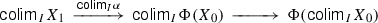For limits, what we have is a diagram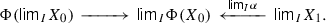(3.3)

To define $$\mathsf{lim}_I X$$, one takes $$(\mathsf{lim}_I X)_0 = \mathsf{lim}_I X_0$$, and one takes the limit $$\Phi (\mathsf{lim}_I X_0) \times _{\mathsf{lim}_I \Phi (X_0)} \mathsf{lim}_I X_1$$ of the diagram (3.3) as $$(\mathsf{lim}_I X)_1$$. $$\square$$

### Proof of Proposition 3.8

For (i), note that $$\langle \mathsf{R}(\Phi ),W \rangle$$ is obviously saturated. Say that a map $$\langle f_0,f_1 \rangle$$ in $$\mathsf{R}(\Phi )$$ is in class F if $$f_0$$ is in F, and the natural map

\begin{aligned} \overline{f}_1:c_1 \rightarrow c_1' \times _{\Phi {c_0'}} \Phi (c_0) \end{aligned}

induced by the commutative square (3.1) is also in F. Since $$\Phi$$ is right-derivable, this is implies that $$f_1$$ is in F, so that it remains to check that C, W, F is a indeed a model structure on $$\mathsf{R}(\Phi )$$.

Definition 2.6 (ii) is obvious, and Definition 2.6 (i) is Lemma 3.9. For Definition 2.6 (iii), assume given a commutative diagram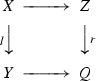in $$\mathsf{R}(\Phi )$$ such that $$l \in C$$, $$r \in F$$, and at least one of the two is in W. To construct a splitting map $$Y \rightarrow Z$$, one first does it for the induced diagram of the components $$X_0,Y_0,Z_0,Q_0 \in \mathcal {C}_0$$. This provides a diagram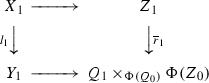(3.4)

in the category $$\mathcal {C}_1$$, and we have to find a splitting map for this diagram. This is indeed possible since by definition, $$l_1$$ is in C, $$\overline{r}_1$$ is in F, and at least one of them is in W.

Finally, for Definition 2.6 (iv), assume given a map $$X \rightarrow Y$$ in $$\mathsf{R}(\Phi )$$. To find either of the two necessary factorizations $$X \rightarrow Z \rightarrow Y$$ for this map, construct first the corresponding factorization $$X_0 \rightarrow Z_0 \rightarrow Y_0$$ in $$\mathcal {C}_0$$, and then factorize the induced map $$X_1 \rightarrow \Phi (Z_0) \times _{\Phi (Y_0)} Y_1$$ in $$\mathcal {C}_1$$.

For (ii), note that since weak equivalences in $$\mathsf{R}(\Phi )$$ are computed componentwise, it suffices to check that a commutative square (2.4) in the homotopy category $${\text {Ho}}(\mathsf{R}(\Phi ),W)$$ is homotopy cartesian resp. cocartesian if and only if so are the corresponding commutative squares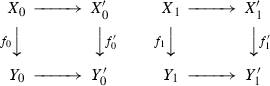(3.5)

in $${\text {Ho}}(\mathcal {C}_0,W_0)$$ and $${\text {Ho}}(\mathcal {C}_1,W_1)$$. Since colimits and cofibrations are also computed componentwise, the cocartesian case is trivial, so let us prove the cartesian case. Again, since weak equivalences are componentwise, we may replace the square with a weakly equivalent one such that $$Y \rightarrow Y'$$ and $$X' \rightarrow Y'$$ are fibrations of fibrant objects, and then by (i), $$Y_0 \rightarrow Y_0'$$, $$X'_0 \rightarrow Y'_0$$, $$Y_1 \rightarrow Y_1'$$ and $$X'_1 \rightarrow Y'_1$$ are also fibrations of fibrant objects. The square (2.4) is then homotopy cocartesian if and only if the map

\begin{aligned} X \rightarrow Y \times _{Y'} X' \end{aligned}

is a weak equivalence, and similarly for the squares (3.5). By the description of the limits in $$\mathsf{R}(\Phi )$$ given in Lemma 3.9, we have $$(Y \times _{Y'} X)_0 \cong Y_0 \times _{Y'_0} X'_0$$, so that to prove the claim, it suffices to prove that the natural map

\begin{aligned} (Y \times _{Y'} X')_1 \rightarrow X_1 \times _{Y'_1} X'_1 \end{aligned}
(3.6)

is a weak equivalence. But since $$\Phi$$ is stable, the natural map

\begin{aligned} \Phi (X_0) \cong \Phi \left( X'_0 \times _{Y_0'} Y_0\right) \rightarrow \Phi (X'_0) \times _{\Phi {Y_0'}} \Phi (Y_0) \end{aligned}

is a weak equivalence, and since $$Y \rightarrow Y'$$, $$X' \rightarrow Y'$$ are fibrations of fibrant objects, the map $$\mathsf{lim}_V \alpha$$ in (3.3) is a fibration. Therefore when we extend the diagram (3.3) to a cartesian square, the square is homotopy cartesian, and (3.6) is indeed a weak equivalence as required. $$\square$$

### Two-sided gluing

Since the notions of a model category and a stable model pair are self-dual, Proposition 3.8 immediately implies an analogous statement for left comma-categories and left-derivable functors. However, it turns out that there is more: one can combine Proposition 3.8 and its dual into a single statement. Namely, assume given two categories $$\mathcal {C}_0$$, $$\mathcal {C}_1$$, two functors $$L,M:\mathcal {C}_0 \rightarrow \mathcal {C}_1$$, and a morphism $$q:L \rightarrow M$$.

### Definition 3.10

The two-sided comma-category $$\mathsf{G}(L,M,q)$$ is the category of quadruples $$\langle c_0,c_1,l,m \rangle$$ of objects $$c_0 \in \mathcal {C}_0$$, $$c_1 \in \mathcal {C}_1$$ equipped with morphisms $$l:L(c_0) \rightarrow c_1$$, $$m:c_1 \rightarrow M(c_0)$$ such that $$m \circ l = q$$.

### Example 3.11

For a real-life example of a two-sided comma-category, consider the standard description of the category of perverse sheaves on a formal disc D, as in e.g. . As $$\mathcal {C}_0$$, we take the category of local systems on the punctured disc $$\overline{D}$$, $$\mathcal {C}_1$$ is the category of sheaves on the central point, $$L=M$$ is the nearby cycles functor $$\Psi$$, and q is identity minus the monodromy operator. Then a perverse sheaf E on the disc corresponds to the quadruple $$\langle E_{\overline{D}},\Phi (E), \mathsf{can}, \mathsf{var}\rangle$$, where $$\Phi$$ is the vanishing cycles functor, and $$\mathsf{can}$$, $$\mathsf{var}$$ are the usual functorial maps.

For any two-sided comma-category of Definition 3.10, forgetting the map m resp. l in a quadruple provides natural functors

\begin{aligned} \lambda ^*:\mathsf{G}(L,M,q) \rightarrow \mathsf{L}(L), \qquad \mu ^*:\mathsf{G}(L,M,q) \rightarrow \mathsf{R}(M) \end{aligned}
(3.7)

to the left resp. right comma-category of the functor L resp. M. We note that if $$\mathcal {C}_1$$ has an initial object 0, and L is the tautological functor sending everything to 0, then $$\mu ^*$$ is an equivalence of categories, so that $$\mathsf{R}(M) \cong \mathsf{G}(L,M,q)$$. Analogously, if $$M(c)=1$$ is a terminal object in $$\mathcal {C}_1$$ for any $$c \in \mathcal {C}_0$$, then $$\mathsf{G}(L,M,q) \cong \mathsf{L}(L)$$.

### Proposition 3.12

1. (i)

Assume that $$\mathcal {C}_0$$, $$\mathcal {C}_1$$ are model categories, L is left-derivable, and M is right-derivable, and equip $$\mathsf{L}(L)$$, $$\mathsf{R}(M)$$ with the model structures of Proposition 3.8. Say that a map f in $$\mathsf{G}(L,M,q)$$ is in C resp. F if so is $$\lambda ^*(f)$$ resp. $$\mu ^*(f)$$, and say that f is in W if $$\lambda ^*(f)$$ is in W (or equivalently, $$\mu ^*(f)$$ is in W). Then C, F, W is a model structure on $$\mathsf{G}(L,M,q)$$.

2. (ii)

Assume given stable model pairs $$\langle \mathcal {C}_0,\mathcal {C}_0'\rangle$$, $$\langle \mathcal {C}_1,\mathcal {C}_1' \rangle$$, assume that the functors L and M are stable in the sense of Definition 3.2, and let $$\mathsf{G}(L,M,q)' \subset \mathsf{G}(L,M,q)$$ be the full subcategory spanned by quadruples $$\langle c_0,c_1,l,m \rangle$$ with $$c_0 \in \mathcal {C}_0'$$, $$c_1 \in \mathcal {C}_1'$$. Then $$\langle \mathsf{G}(L,M,q)', \mathsf{G}(L,M,q) \rangle$$ is a stable model pair.

### Proof

For (i), note that a map in $$\mathsf{G}(L,M,q)$$ is a weak equivalence iff it is a weak equivalence componentwise, so that $$\mathsf{G}(L,M,q)$$ is trivially saturated, and moreover, Definition 2.6 (ii) tautologically follows from the corresponding property of the model structures on $$\mathsf{L}(L)$$ and $$\mathsf{R}(M)$$.

To check Definition 2.6 (i), assume given a finite category I and a functor $$X:I \rightarrow \mathsf{G}(L,M,q)$$, set $$(\mathsf{lim}_I X)_0 = \mathsf{lim}X_0$$, define $$(\mathsf{lim}_I X)_1$$ and a map $$\widetilde{m}$$ by the cartesian diagram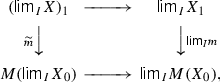and define a map $$\widetilde{l}:L(\mathsf{lim}_I X_0) \rightarrow (\mathsf{lim}_I X)_1$$ as the product of the map q and the composition map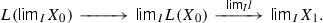Then $$\langle (\mathsf{lim}_I X)_0,(\mathsf{lim}_I X)_1,\widetilde{l},\widetilde{m} \rangle$$ is a well-defined object in $$\mathsf{G}(L,M,q)$$, and it is easy to see that it gives the limit of the functor X. Therefore $$\mathsf{G}(L,M,q)$$ has finite limits, and moreover, $$\mu ^*$$ preserves then. Analogously, $$\mathsf{G}(L,M,q)$$ has finite colimits, and $$\lambda ^*$$ preserves them.

For Definition 2.6 (iii), the argument is the same as in the proof of Proposition 3.8, except that in the second step, instead of splitting the diagram (3.4), one splits a natural diagram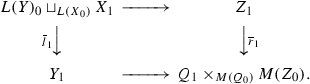For Definition 2.6 (iv), the argument is again essentially the same as in Proposition 3.8: to find a factorization $$X \rightarrow Z \rightarrow Y$$ of a map $$X \rightarrow Y$$, one first finds a factorization $$X_0 \rightarrow Z_0 \rightarrow Y_0$$ of the corresponding map $$X_0 \rightarrow Y_0$$, and the factorizes the induced natural map

\begin{aligned} L(Z_0) \sqcup _{L(X_0)} X_1 \rightarrow Y_1 \times _{M(Y_0)} M(Z_0). \end{aligned}

This finishes the proof of (i).

For (ii), observe that again as in Proposition 3.8 it suffices to prove that a square (2.4) is homotopy cartesian resp. cocartesian if and only if so are the squares (3.5). In the cartesian case, note that $$\mu ^*$$ sends weak equivalence to weak equivalences, fibrations to fibrations, and cartesian squares to cartesian squares, so that (2.4) is homotopy cartesian iff it becomes homotopy cartesian after applying the functor $$\mu ^*$$. The claim then immediately follows from the corresponding claim in the proof of Proposition 3.8. In the cocartesian case, use the functor $$\lambda ^*$$. $$\square$$

### Semiorthogonal decompositions

To understand better what are the implications of Proposition 3.12 (ii) on the level of homotopy categories, let us briefly recall the Verdier localization formalism and the notion of a semiorthogonal decomposition.

A full triangulated subcategory $${\mathcal {D}}_0 \subset {\mathcal {D}}$$ in a triangulated category $${\mathcal {D}}$$ is called thick, or sometimes saturated, if it is closed under retracts ( has a formally different notion of an “epaisse” subcategory that turns out to be equivalent).

### Theorem 3.13

() Assume given a thick full triangulated subcategory $${\mathcal {D}}_0 \subset {\mathcal {D}}$$ in a triangulated category $${\mathcal {D}}$$.

1. (i)

The class W of maps f in $${\mathcal {D}}$$ whose cone $${\text {Cone}}(f)$$ lies in $${\mathcal {D}}_0$$ is saturated in the sense of Definition 2.2.

2. (ii)

Assume that the relative category $$\langle {\mathcal {D}},W \rangle$$ is localizable in the sense of Definition 2.4. Then the homotopy category $${\text {Ho}}({\mathcal {D}},W)$$ is triangulated, the quotient functor $$h:{\mathcal {D}}\rightarrow {\text {Ho}}({\mathcal {D}},W)$$ is a triangulated functor, and for any object $$E \in {\mathcal {D}}$$, $$h(E)=0$$ if and only if E lies in $${\mathcal {D}}_0 \subset {\mathcal {D}}$$. $$\square$$

When the condition (ii) of Theorem 3.13 is satisfied, so that $${\text {Ho}}({\mathcal {D}},W)$$ exists, one usually calls it the quotient category of $${\mathcal {D}}$$ by $${\mathcal {D}}_0$$ and denotes it by $${\mathcal {D}}/{\mathcal {D}}_0$$. This condition is not in , and in fact Verdier himself did not split his theorem into two parts. He did not need to: he was working in Grothendieck’s set theory setup with the universe axiom, and in this setup, any relative category is trivially localizable, although the quotient $${\mathcal {D}}/{\mathcal {D}}_0$$ might belong to a bigger universe. If one does not assume the freedom to enlarge universes at will, one has to somehow control the size of the quotient. What Verdier showed is that morphisms in $${\mathcal {D}}/{\mathcal {D}}_0$$ can always be represented by diagrams (2.1) of length at most 2, in either of the two ways possible depending on your preference ($$w^{-1} \circ f$$ or $$f \circ w^{-1}$$). Then if for any object $$E \in {\mathcal {D}}$$, one denotes by W(E) the category of objects $$E' \in {\mathcal {D}}$$ equipped with a map $$w:E \rightarrow E'$$ in the class W, the resulting category W(E) is filtered, and morphisms in the quotient category $${\mathcal {D}}/{\mathcal {D}}_0$$ are given by

\begin{aligned} {\text {Hom}}(h(A),h(B)) = \mathsf{colim}_{B' \in W(B)}{\text {Hom}}(A,B'). \end{aligned}
(3.8)

One has to insure that the colimit makes sense. For example, one can do it by imposing the following condition.

### Definition 3.14

A full triangulated subcategory $${\mathcal {D}}_0 \subset {\mathcal {D}}$$ in a triangulated category $${\mathcal {D}}$$ is right-localizing if for any $$E \in {\mathcal {D}}$$, there exists a filtered small category I and a cofinal functor $$I \rightarrow W(E)$$, and left-localizing if $${\mathcal {D}}^o_0 \subset {\mathcal {D}}^o$$ is right-localizing.

If $${\mathcal {D}}_0 \subset {\mathcal {D}}$$ is right-localizing, then (3.8) gives a well-defined set, so that $$\langle {\mathcal {D}},W \rangle$$ is localizable, and the quotient $${\mathcal {D}}/{\mathcal {D}}_0$$ exists. Dually, if $${\mathcal {D}}_0 \subset {\mathcal {D}}$$ is left-localizing, the quotient $${\mathcal {D}}/{\mathcal {D}}_0 = ({\mathcal {D}}^o/{\mathcal {D}}_0^o)^o$$ also exists. A small subcategory $${\mathcal {D}}_0 \subset {\mathcal {D}}$$ is always both right and left-localizing: up to an isomorphism, an object $$w:E' \rightarrow E$$ in W(E) is given by an object $${\text {Cone}}(w) \in {\mathcal {D}}_0$$ and a morphism $$E \rightarrow {\text {Cone}}(w)$$, so that W(E) itself is essentially small. Another example of a localizing triangulated subcategory appears in the situation of a semiorthogonal decomposition introduced in .

### Definition 3.15

A semiorthogonal decomposition $$\langle {\mathcal {D}}_0,{\mathcal {D}}_1 \rangle$$ of a triangulated category $${\mathcal {D}}$$ consists of two full triangulated subcategories $${\mathcal {D}}_0,{\mathcal {D}}_1 \subset {\mathcal {D}}$$ such that

1. (i)

$${\text {Hom}}(A,B) = 0$$ for any $$A \in {\mathcal {D}}_0 \subset {\mathcal {D}}$$, $$B \in {\mathcal {D}}_1 \subset {\mathcal {D}}$$, and

2. (ii)

any object $$E \in {\mathcal {D}}$$ fits into a distinguished triangle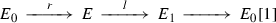(3.9)

in $${\mathcal {D}}$$ with $$E_0 \in {\mathcal {D}}_0$$ and $$E_1 \in {\mathcal {D}}_1$$.

One shows that in the situation of Definition 3.15, $$E_0$$ and $$E_1$$ in (3.9) are functorial in E, and sending E to $$E_0$$ resp. $$E_1$$ gives functors $$R:{\mathcal {D}}\rightarrow {\mathcal {D}}_0$$, $$L:{\mathcal {D}}\rightarrow {\mathcal {D}}_1$$ right resp. left-adjoint to the embeddings, with r and l of (3.9) being the adjunction map. Both $${\mathcal {D}}_0$$ and $${\mathcal {D}}_1$$ are automatically thick, $${\mathcal {D}}_0 \subset {\mathcal {D}}$$ is right-localizing, $${\mathcal {D}}_1 \subset {\mathcal {D}}$$ is left-localizing, and L, R induce equivalences $${\mathcal {D}}_1 \cong {\mathcal {D}}/{\mathcal {D}}_0$$, $${\mathcal {D}}_0 \cong {\mathcal {D}}/{\mathcal {D}}_1$$.

Conversely, a full triangulated subcategory $${\mathcal {D}}_0 \subset {\mathcal {D}}_1$$ is called left resp. right-admissible if the embedding functor admits a left resp. right-adjoint, and simply admissible if it is left and right-admissible. Then any right-admissible full triangulated subcategory $${\mathcal {D}}_0 \subset {\mathcal {D}}$$ is automatically thick and right-localizing, the quotient functor $${\mathcal {D}}\rightarrow {\mathcal {D}}_1 = {\mathcal {D}}/{\mathcal {D}}_0$$ has a fully faithful right-adjoint $${\mathcal {D}}_1 \rightarrow {\mathcal {D}}$$, and $$\langle {\mathcal {D}}_0,{\mathcal {D}}_1 \rangle$$ is a semiorthogonal decomposition of the category $${\mathcal {D}}$$. Dually, for a left-admissible subcategory $${\mathcal {D}}_0 \subset {\mathcal {D}}$$, we have a left-adjoint $${\mathcal {D}}_1 = {\mathcal {D}}/{\mathcal {D}}_0 \rightarrow {\mathcal {D}}$$ to the quotient functor, and a semiorthogonal decomposition $$\langle {\mathcal {D}}_1,{\mathcal {D}}_0 \rangle$$.

For an admissible subcategory $${\mathcal {D}}_0 \subset {\mathcal {D}}$$, we can actually compose the embedding $${\mathcal {D}}/{\mathcal {D}}_0 \rightarrow {\mathcal {D}}$$ right-adjoint to the quotient functor with the left-adjoint $${\mathcal {D}}\rightarrow {\mathcal {D}}_0$$ to the embedding, and obtain a triangulated functor $$F:{\mathcal {D}}/{\mathcal {D}}_0 \rightarrow {\mathcal {D}}_0$$. This is known as the left gluing functor of the semiorthogonal decomposition $$\langle {\mathcal {D}}_0,{\mathcal {D}}/{\mathcal {D}}_0 \rangle$$. Alternatively, we can take the left-adjoint $${\mathcal {D}}/{\mathcal {D}}_0 \rightarrow {\mathcal {D}}$$ and the right-adjoint $${\mathcal {D}}\rightarrow {\mathcal {D}}_0$$; this gives the right gluing functor $$F':{\mathcal {D}}/{\mathcal {D}}_0 \rightarrow {\mathcal {D}}_0$$.

Now, doing a version of Theorem 3.13 for stable model pairs is rather delicate, and we will do it in the end of the paper, in Sect. 12. However, in the situation of a semiorthogonal decomposition, things are straightforward. Namely, if we have a stable model pair $$\langle \mathcal {C},\mathcal {C}' \rangle$$ and a semiorthogonal decomposition $$\langle {\mathcal {D}}_0,{\mathcal {D}}_1 \rangle$$ of the triangulated category $${\mathcal {D}}= {\text {Ho}}(\mathcal {C})$$, then the preimages $$\mathcal {C}_0,\mathcal {C}_1 \subset \mathcal {C}$$ of the subcategories $${\mathcal {D}}_0,{\mathcal {D}}_1 \subset {\mathcal {D}}$$ under the localization functor $$h:\mathcal {C}\rightarrow {\mathcal {D}}$$ trivially fit into stable model pairs $$\langle \mathcal {C}_0,\mathcal {C}' \rangle$$, $$\langle \mathcal {C}_1,\mathcal {C}' \rangle$$.

Let us now show that in a sense, Proposition 3.12 (ii) reverses the construction and produces a triangulated category starting from gluing data for a semiorthogonal decomposition.

In the setup of Proposition 3.12 (ii), the category $${\text {Ho}}(\mathsf{G}(L,M,q)')$$ is triangulated by Proposition 2.20, and so are the categories $${\text {Ho}}(\mathcal {C}_0')$$, $${\text {Ho}}(\mathcal {C}_1')$$. Denote by $$U:\mathsf{G}(L,M,q') \rightarrow \mathcal {C}_0$$ the natural forgetful functor sending $$\langle X_0,X_1,l,m \rangle$$ to $$X_0$$, and note that setting

\begin{aligned} U_\dagger (X) = \langle X,L(X),\mathsf{id},q \rangle , \quad U^\dagger (X) = \langle X,M(X),q,\mathsf{id}\rangle , \qquad X \in \mathcal {C}_0' \end{aligned}

defines functors $$U_\dagger ,U^\dagger :\mathcal {C}_0 \rightarrow \mathsf{G}(L,M,q)$$ left resp. right-adjoint to $$\pi$$. Moreover, let

\begin{aligned} V:\mathcal {C}_1 \rightarrow \mathsf{G}(L,M,q) \end{aligned}
(3.10)

be the functor sending $$X \in \mathcal {C}_1$$ to $$\langle 0,X,0,0 \rangle$$, and note that it has a left resp. right-adjoint functors $$V_\dagger$$, $$V^\dagger$$ defined by the cocartesian resp. cartesian square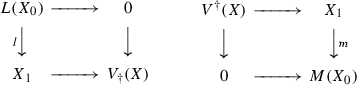(3.11)

in $$\mathcal {C}_1$$ for any $$X = \langle X_0,X_1,l,m \rangle \in \mathsf{G}(L,M,q)$$.

### Lemma 3.16

In the situation of Proposition 3.8 (ii), the functors U and V are left and right-derivable, the functors $$U_\dagger$$ and $$V_\dagger$$ are left-derivable, and the functors $$U^\dagger$$ and $$V^\dagger$$ are right-derivable. All these functors are stable in the sense of Definition 3.2. The derived functor $$L^\cdot V \cong R^\cdot V$$ is fully faithful, and $$L^\cdot V_\dagger$$ resp. $$R^\cdot V^\dagger$$ is adjoint to it on the left and on the right. For any object $$X = \langle X_0,X_1,l,m \rangle \in {\text {Ho}}(\mathsf{G}(L,M,q)')$$, we have functorial distinguished triangles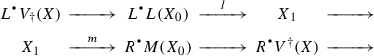(3.12)

in the triangulated category $${\text {Ho}}(\mathcal {C}_1')$$. The derived functors $$L^\cdot U_\dagger$$ resp. $$R^\cdot U^\dagger$$ are both fully faithful and left resp. right-adjoint to $$R^\cdot U \cong L^\cdot U$$, so that we have semiorthogonal decompositions

\begin{aligned} \langle V({\text {Ho}}(\mathcal {C}_1)),R^\cdot U^\dagger ({\text {Ho}}(\mathcal {C}_0)) \rangle , \qquad \langle L^\cdot U_\dagger ({\text {Ho}}(\mathcal {C}_0)),V({\text {Ho}}(\mathcal {C}_1)) \rangle \end{aligned}

of the category $${\text {Ho}}(\mathsf{G}(L,M,q)')$$ in the sense of Definition 3.15.

### Proof

The fact that U and V are both left and right-derivable follows immediately from the definition of the model structure on $$\mathsf{G}(L',M',q')$$. Then by adjunction, $$U_\dagger$$ and $$V_\dagger$$ resp. $$U^\dagger$$ and $$V^\dagger$$ are left resp. right-derivable. Moreover, all these functors are in fact Quillen, so that they are all automatically stable, and we obtain the required adjunctions on the homotopy category level by the Quillen Adjunction Theorem. The functor V is also obviously fully faithful, and we have $$R^\cdot V^\dagger \circ R^\cdot V \cong R^\cdot (V^\dagger \circ V) \cong \mathsf{id}$$, so that $$L^\cdot V \cong R^\cdot V$$ is still fully faithful. The distinguished triangles (3.12) are induced by the squares (3.11). Finally, the functors $$U_\dagger$$ and $$U^\dagger$$ are also obviously fully faithful, and since U is both left and right-derivable, their derived functors are fully faithful as well. $$\square$$

## Families of categories

### Grothendieck construction

From the point of view of category theory, the right and left comma-categories are the most elementary examples of gluing. To see what happens in more complicated situations, let us try to iterate the construction. Namely, assume given two categories $$\mathcal {C}_1$$, $$\mathcal {C}_2$$ with a functor $$\Phi _{12}:\mathcal {C}_1 \rightarrow \mathcal {C}_2$$, consider the right comma-category $$\mathsf{R}(\Phi _{12})$$, and assume given a third category $$\mathcal {C}_0$$. Then by definition, a functor $$\Phi :\mathcal {C}_0 \rightarrow \mathsf{R}(\Phi _{12})$$ is given by a triple $$\langle \Phi _{01},\Phi _{02},\alpha \rangle$$ of two functors $$\Phi _{01}:\mathcal {C}_0 \rightarrow \mathcal {C}_1$$, $$\Phi _{01}:\mathcal {C}_0 \rightarrow \mathcal {C}_2$$, and a morphism

\begin{aligned} \alpha _{012}:\Phi _{02} \rightarrow \Phi _{12} \circ \Phi _{01}. \end{aligned}
(4.1)

The comma-category $$\mathsf{R}(\Phi )$$ of such a functor is then the category of triples $$\langle c_0,c_1,c_2 \rangle$$ of objects $$c_l \in \mathcal {C}_l$$, $$l=0,1,2$$, equipped with morphisms $$\alpha _{01}:c_1 \rightarrow \Phi _{01}(c_0)$$, $$\alpha _{02}:c_2 \rightarrow \Phi _{02}(c_0)$$, $$\alpha _{12}:c_2 \rightarrow \Phi _{12}(c_1)$$ such that $$\Phi _{12}(\alpha _{01}) \circ \alpha _{12} = \alpha _{012} \circ \alpha _{02}$$. More generally, doing the procedure n times, we end up with categories $$\mathcal {C}_i$$, $$0 \le i \le n$$ connected by functors $$\Phi _{ij}:\mathcal {C}_i \rightarrow \mathcal {C}_j$$, $$1 \le i < j \le n$$, and maps $$\alpha _{ijk}:\Phi _{ik} \rightarrow \Phi _{jk} \circ \Phi _{ij}$$, $$0 \le i< j < k \le n$$, such that $$\alpha _{ijk} \circ \alpha _{ikl} = \alpha _{jkl} \circ \alpha _{ijl}$$, $$0 \le i< j< k < l \le n$$. A convenient way to package data of this kind is the formalism of . We summarize it as follows.

### Definition 4.1

A morphism $$f:c' \rightarrow c$$ in a category $$\mathcal {C}$$ is vertical with respect to a functor $$\pi :\mathcal {C}\rightarrow I$$ if $$\pi (f)=\mathsf{id}$$, and cartesian with respect to $$\pi$$ if any $$f':c'' \rightarrow c$$ such that $$\pi (f) = \pi (f')$$ uniquely factorizes as $$f' = f \circ f_0$$ with vertical $$f_0$$. A cartesian lifting of a morphism $$f:i' \rightarrow i$$ in I is a morphism $$f'$$ in $$\mathcal {C}$$ cartesian with respect to $$\pi$$ and such that $$\pi (f')=f$$. A functor $$\pi :\mathcal {C}\rightarrow I$$ is a prefibration if for any $$c \in \mathcal {C}$$, any morphism $$f:i' \rightarrow i=\pi (c)$$ in $$\mathcal {E}$$ admits a cartesian lifting $$f':c' \rightarrow c$$. A prefibration is a fibration if the composition of cartesian morphisms is cartesian. A morphism is cocartesian if it is cartesian as a morphism in the opposite category $$\mathcal {C}^o$$ with respect to the opposite functor $$\pi ^o:\mathcal {C}^o \rightarrow I^o$$, and a functor $$\pi$$ is a precofibration resp. cofibration if the opposite functor $$\pi ^o$$ is a prefibration resp. fibration. A functor $$\pi$$ is a bifibration if it is both a fibration and a cofibration.

### Remark 4.2

In recent retellings of  such as , it has become usual to strengthen the definition of a cartesian map so that every prefibration is automatically a fibration. However, for our purposes, prefibrations are just as important.

### Remark 4.3

The reader will immediately notice that the terminology of Definition 4.1 clashes with the standard terminology for model categories. Unfortunately, there is no easy way out of this, since both are well-established. Fortunately, we will mostly need prefibrations and precofibrations of Definition 4.1, not fibrations and cofibrations. To handle the remaining possible conflicts, from now on, we adopt the following convention:

1. (i)

In Sects. 4, 5 and 6, model structures do not appear at all. In these Sections, “fibration” resp. “cofibration” means fibration resp. cofibration in the sense of Definition 4.1.

2. (ii)

From Sect. 7 onward, “fibration” or “cofibration” without qualifiers is always used in the model category sense, and fibrations resp. cofibrations of Definition 4.1 are Grothendieck fibrations resp. Grothendieck cofibrations.

### Example 4.4

For any functor $$\Phi :\mathcal {C}_0 \rightarrow \mathcal {C}_1$$ between two categories $$\mathcal {C}_0$$, $$\mathcal {C}_1$$, the projection $$\tau :\mathsf{R}(\Phi ) \rightarrow \mathcal {C}_1$$ of (3.2) is a fibration, and dually, the projection $$\tau :\mathsf{L}(\Phi ) \rightarrow \mathcal {C}_1$$ is a cofibration. A map f is cartesian resp. cocartesian with respect to $$\tau$$ iff $$\sigma (f)$$ is invertible.

If $$\pi :\mathcal {C}\rightarrow I$$ is a prefibration, then cartesian liftings of morphisms in I are defined by their targets up to a canonical isomorphism, and sending $$c \in \mathcal {C}$$ to the source $$c'$$ of the cartesian lifting $$f'$$ of a morphism $$f:i' \rightarrow i=\pi (c)$$ gives a functor $$f^*:\mathcal {C}_{i} \rightarrow \mathcal {C}_{i'}$$ between the fibers $$\mathcal {C}_{i} = \pi ^{-1}(i)$$, $$\mathcal {C}_{i'} = \pi ^{-1}(i')$$ of the fibration $$\pi$$. These are called the transition functors of the prefibration. For an identity map $$\mathsf{id}:i \rightarrow i$$ in I, we have $$\mathsf{id}^*=\mathsf{id}$$. For any pair $$f_1$$, $$f_2$$ of composable morphisms, we have a natural map

\begin{aligned} \alpha (f_1,f_2):f_2^* \circ f_1^* \rightarrow (f_1 \circ f_2)^*, \end{aligned}
(4.2)

we have $$\alpha (\mathsf{id},f)=\alpha (f,\mathsf{id})=\mathsf{id}$$, and and for any triple $$f_1$$, $$f_2$$, $$f_3$$ of composable morphisms, the square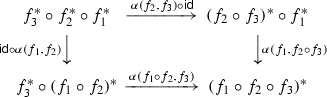(4.3)

is commutative. Axiomatizing the situation, one arrives at the following.

### Definition 4.5

A covariant lax functor F from a category I to the 2-category $${\text {Cat}}$$ of categories is a collection of the following data:

1. (i)

a category F(i) for any object $$i \in I$$,

2. (ii)

a functor $$F(f):F(i) \rightarrow F(i')$$ for any morphism $$f:i \rightarrow i'$$ in I,

3. (iii)

a morphism $$\alpha (i):F(\mathsf{id}_i) \rightarrow \mathsf{id}_{F(i)}$$ for any identity map $$\mathsf{id}_i:i \rightarrow i$$, and

4. (iv)

a morphism $$\alpha (f_1,f_2):F(f_1 \circ f_2) \rightarrow F(f_1) \circ F(f_2)$$ for any composable pair of maps $$f_1$$, $$f_2$$,

such that $$(\alpha (i) \circ \mathsf{id}) \circ \alpha (\mathsf{id}_i,f) = \mathsf{id}$$, $$(\mathsf{id}\circ \alpha (i')) \circ \alpha (f,\mathsf{id}_{i'})=\mathsf{id}$$ for any $$f:i \rightarrow i'$$, and $$(\alpha (f_1 \circ f_2) \circ \mathsf{id}) \circ \alpha (f_1 \circ f_2,f_3) = (\mathsf{id}\circ \alpha (f_2,f_3)) \circ \alpha (f_1,f_2 \circ f_3)$$ for any composable triple $$f_1$$, $$f_2$$, $$f_3$$. A lax functor is normalized if $$\alpha (i)$$ is an isomorphism for any $$i \in I$$. A contravariant lax functor from I to $${\text {Cat}}$$ is a covariant lax functor from $$I^o$$ to $${\text {Cat}}$$.

Then in this terminology, a prefibration I defines a normalized contravariant lax functor from I to $${\text {Cat}}$$.

A prefibration is a fibration if and only if all the maps (4.2) are invertible. In this case, the corresponding lax functor reduces to what Grothendieck called a contravariant pseudofunctor from I to $${\text {Cat}}$$. Conversely, for any such pseudofunctor $$F:I^o \rightarrow {\text {Cat}}$$, one can consider the category $$\mathcal {C}$$ of pairs $$\langle i,c \rangle$$, $$i \in I$$, $$c \in F(i)$$, with maps from $$\langle i,c \rangle$$ to $$\langle i',c' \rangle$$ given by a map $$f:i \rightarrow i'$$ and a map $$c \rightarrow F(f)(c')$$, and composition defined using the morphisms $$\alpha (f,f')$$. The two constructions are inverse to each other (nowadays this is known as the Grothendieck construction). The same inverse construction identifies prefibrations and normalized lax functors.

By a dual construction, cofibrations $$\mathcal {C}\rightarrow I$$ correspond to covariant pseudofunctors, with transition functors $$f_!:\mathcal {C}_i \rightarrow \mathcal {C}_{i'}$$ corresponding to maps $$f:i \rightarrow i'$$, and natural isomorphisms

\begin{aligned} \alpha (f_1,f_2):(f_1 \circ f_2)_! \rightarrow f_{1!} \circ f_{2!} \end{aligned}
(4.4)

for any pair $$f_1$$, $$f_2$$ of composable maps. For a precofibration, the maps (4.4) do not have to be isomorphisms, and we only get a normalized covariant lax functor.

### Remark 4.6

Strictly speaking, transition functors $$f^*$$ of a prefibration are well-defined only up to a unique isomorphism, not uniquely. To convert a prefibration into a lax functor, one has to actually fix the functors $$f^*$$ (in Grothendieck’s terminology, this is called choosing a cleavage of a prefibration). However, lax functors obtained from different cleavages are canonically isomorphic.

### Example 4.7

Let $$\Delta$$ be the category of finite ordinals $$[n] = \{0,\dots ,n\}$$, $$n \ge 0$$ and order-preserving maps between them. Note that we can treat an ordinal as a small category in the usual way, and then this is firstly, consistent with our earlier notation, and secondly, gives an embedding $$\Delta \subset {\text {Cat}}$$. The Grothendieck construction then associates a cofibration $$\nu :\Delta ^\flat \rightarrow \Delta$$ to this embedding. Objects of the category $$\Delta ^\flat$$ are pairs $$\langle [n],l \rangle$$, $$[n] \in \Delta$$, $$l \in [n]$$, and maps from $$\langle [n],l \rangle$$ to $$\langle [n'],l' \rangle$$ given by maps $$f:[n] \rightarrow [n']$$ such that $$f(l) \le l'$$. The functor $$\nu$$ is the forgetful functor sending $$\langle [n],l \rangle$$ to [n].

Alternatively, one can send $$[n] \in \Delta$$ to the opposite category $$[n]^o$$. This gives the category $$\Delta _\flat$$ of pairs $$\langle [n],l \rangle$$ and maps $$f:[n] \rightarrow [n']$$ such that $$f(l) \ge l'$$, again with the forgetful functor $$\nu :\Delta _\flat \rightarrow \Delta$$.

### Remark 4.8

The category $$\Delta$$ is embedded into the larger category of all finite non-empty totally ordered sets, and although the embedding is an equivalence, it is prudent to distinguish between the two (as e.g. in ). We will sometimes fail to do so and assume that, by abuse of notation, any abstract finite non-empty ordinal defines an object in $$\Delta$$. Doing this consistently amounts to fixing once and for all an equivalence inverse to the embedding above, and it is certainly possible to do this in any reasonable foundational context.

### Example 4.9

A useful source of prefibrations is the following construction. Assume given a prefibration $$\mathcal {C}\rightarrow I$$ and a full subcategory $$\mathcal {C}' \rightarrow I$$. Moreover, assume that either for any object $$i \in I$$, the embedding $$\mathcal {C}'_i \rightarrow \mathcal {C}_i$$ admits a right-adjoint functor r(i), or for any morphism $$f:i \rightarrow i'$$, the transition functor $$f^*:\mathcal {C}_{i'} \rightarrow \mathcal {C}_i$$ sends $$\mathcal {C}'_{i'} \subset \mathcal {C}_{i'}$$ into $$\mathcal {C}'_i \subset \mathcal {C}_i$$. Then the induced functor $$\mathcal {C}' \rightarrow I$$ is also a prefibration. In the second case, its transition functors are simply induced by the transition functors $$f^*$$ of $$\mathcal {C}\rightarrow I$$, and in the first case, they are given by $$r(i) \circ f^*$$ for any $$f:i \rightarrow i'$$.

### Functors and sections

Assume given categories $$\mathcal {C}$$, $$\mathcal {C}'$$ and functors $$\pi :\mathcal {C}\rightarrow I$$, $$\pi ':\mathcal {C}' \rightarrow I$$ to a category I.

### Definition 4.10

1. (i)

A functor from $$\mathcal {C}$$ to $$\mathcal {C}'$$ over I is a functor $$F:\mathcal {C}\rightarrow \mathcal {C}'$$ equipped with an isomorphism $$\pi ' \circ F \cong \pi$$.

2. (ii)

Assume that $$\pi$$, $$\pi '$$ are prefibrations. A functor F from $$\mathcal {C}$$ to $$\mathcal {C}'$$ is cartesian along a morphism $$f:i \rightarrow i'$$ in I if it sends cartesian liftings of f to cartesian liftings of f. A functor F is cartesian if it sends all cartesian maps to cartesian maps.

Explicitly, for any two categories $$\mathcal {C}$$, $$\mathcal {C}'$$ equipped with prefibrations over I, a functor $$F:\mathcal {C}\rightarrow \mathcal {C}'$$ over I defines functors $$F(i):\mathcal {C}_i \rightarrow \mathcal {C}'_i$$ for any $$i \in I$$ and morphisms

\begin{aligned} F(f):F(i) \circ f^* \rightarrow f^* \circ F(i') \end{aligned}
(4.5)

for any morphism $$f:i \rightarrow i'$$ in I compatible with the maps (4.2) (we leave it to an interested reader to write down the explicit compatibility condition). The functor F is cartesian over f iff the morphism (4.5) is an isomorphism.

### Lemma 4.11

Assume given two prefibrations $$\pi :\mathcal {C}\rightarrow I'$$, $$\gamma :I' \rightarrow I$$. Then the following conditions are equivalent.

1. (i)

The composition functor $$\gamma \circ \pi :\mathcal {C}\rightarrow I$$ is a prefibration, and $$\pi$$ is cartesian over I.

2. (ii)

The map (4.2) for the prefibration $$\pi$$ is an isomorphism as soon as $$f_1$$ is cartesian and $$f_2$$ is vertical over I.

### Proof

It immediately follows from the definition that any map f in $$\mathcal {C}$$ cartesian over I must be also cartesian over $$I'$$. Therefore (i) is equivalent to saying that any map f in $$\mathcal {C}$$ cartesian over $$I'$$ and such that $$\pi (f)$$ is cartesian over I is itself cartesian over I. Let $$f:c' \rightarrow c$$ be such a map. Then since $$\pi$$ is a prefibration, any other map $$f':c'' \rightarrow c$$ in $$\mathcal {C}$$ must factor uniquely as $$f' = f'' \circ v$$ with $$f''$$ cartesian and v vertical over $$I'$$, and if $$\gamma (\pi (f)) = \gamma (\pi (f'))$$, then we must also have $$\pi (f') = \pi (f) \circ v'$$, where $$v':\pi (c'') \rightarrow \pi (c')$$ is vertical over I. Now, if we denote by $$v'':v^*c' \rightarrow c'$$ the cartesian lifting of the map $$v'$$, then the cartesian property of f over I is equivalent to saying that $$f'$$ factors uniquely through $$f \circ v''$$ by means of a map vertical over $$I'$$, so that $$f \circ v''$$ has the same universal property as $$f''$$. Then we must have $$f \circ v'' = f''$$, and this is equivalent to (ii). $$\square$$

### Lemma 4.12

Assume given a prefibration $$\pi :\mathcal {C}\rightarrow I'$$ and a precofibration $$\gamma :I' \rightarrow I$$. Then the following conditions are equivalent.

1. (i)

The composition functor $$\gamma \circ \pi :\mathcal {C}\rightarrow I$$ is a precofibration, and $$\pi$$ is cocartesian over I.

2. (ii)

The map (4.2) for the prefibration $$\pi$$ is an isomorphism as soon as $$f_2$$ is cocartesian and $$f_1$$ is vertical over I, and for any map f in $$I'$$ cocartesian over I, the transition functor $$f^*$$ admits a left-adjoint $$f_!$$.

### Proof

By definition, (i) is equivalent to saying that for any $$c \in \mathcal {C}$$ and map $$f:\pi (c) \rightarrow i'$$ cocartesian over I, there exists a map $$f':c \rightarrow c'$$ that lifts f (that is, $$\pi (f')=f$$), and is cocartesian both over $$I'$$ and over I. Existence of lifting maps $$f'$$ cocartesian over $$I'$$ is equivalent to saying that $$f^*$$ has a left-adjoint $$f_!$$. As in Lemma 4.2, the condition on the maps (4.2) is then equivalent to saying that these maps $$f'$$ are also cocartesian over I. $$\square$$

A section of a prefibration $$\pi :\mathcal {C}\rightarrow I$$ is a functor $$\sigma :I \rightarrow \mathcal {C}$$ equipped with an isomorphism $$\pi \circ \sigma \cong \mathsf{id}$$. Equivalently, $$\sigma$$ is a functor from I to $$\mathcal {C}$$ over I in the sense of Definition 4.10 (i), where I is equipped with the identity functor $$\mathsf{id}:I \rightarrow I$$. A section is explicitly given by specifying a collection of objects $$\sigma (i) \in \mathcal {C}_i$$, $$i \in I$$, and a map

\begin{aligned} \sigma (f):\sigma (i') \rightarrow f^*\sigma (i) \end{aligned}
(4.6)

for any morphism $$f:i' \rightarrow i$$ in I, subject to compatibility conditions. If I is small, then sections of a prefibration $$\mathcal {C}\rightarrow I$$ form a category that we denote by $${\text {Sec}}(I,\mathcal {C})$$, and sending a section $$\sigma$$ to the object $$\sigma (i)$$ gives a functor

\begin{aligned} \mathsf{ev}_i:{\text {Sec}}(I,\mathcal {C}) \rightarrow \mathcal {C}_i \end{aligned}
(4.7)

that we call “evaluation at i”. A section $$\sigma$$ is cartesian along a morphism f if it is cartesian as a functor (equivalently, $$\sigma (f)$$ is cartesian over I, equivalently, (4.6) is an isomorphism). We denote by

\begin{aligned} {\text {Sec}}_\natural (I,\mathcal {C}) \subset {\text {Sec}}(I,\mathcal {C}) \end{aligned}
(4.8)

the full subcategory spanned by sections cartesian along all morphisms in I.

Dually, a functor $$F:\mathcal {C}_1 \rightarrow \mathcal {C}_2$$ between two precofibrations is cocartesian along a morphism f if $$F^o$$ is cartesian along f, and for any cofibration $$\mathcal {C}\rightarrow I$$ over a small category I, we denote by

\begin{aligned} {\text {Sec}}^\natural (I,\mathcal {C}) \subset {\text {Sec}}(I,\mathcal {C}) \end{aligned}
(4.9)

the full subcategory spanned by cocartesian sections. Note that if $$\mathcal {C}\rightarrow I$$ is a bifibration, then both $${\text {Sec}}_\natural (I,\mathcal {C})$$ and $${\text {Sec}}^\natural (I,\mathcal {C})$$ are well-defined; in general, these categories are completely different.

### Example 4.13

Let $$I=^o$$. By definition, a prefibration $$\mathcal {C}$$ over I consists of two categories $$\mathcal {C}_0$$, $$\mathcal {C}_1$$, and a transition functor $$\Phi :\mathcal {C}_0 \rightarrow \mathcal {C}_1$$. The category $${\text {Sec}}(^o,\mathcal {C})$$ is then precisely the right comma-category $$\mathsf{R}(\Phi )$$, and the evaluation functors $$\mathsf{ev}_0$$ resp. $$\mathsf{ev}_1$$ send $$\langle c_0,c_1,\alpha \rangle$$ to $$c_0$$ resp. $$c_1$$.

### Example 4.14

In the situation of Example 4.13, assume that $$\mathcal {C}_0$$ and $$\mathcal {C}_1$$ are equipped with prefibrations $$\mathcal {C}_0,\mathcal {C}_1 \rightarrow I$$, and $$\Phi$$ is a functor over I. Then $$\mathcal {C}\rightarrow I \times ^o$$ is also a prefibration, and we have $${\text {Sec}}(I \times ,\mathcal {C}) \cong \mathsf{R}({\text {Sec}}(I,\Phi ))$$, where $${\text {Sec}}(I,\Phi ):{\text {Sec}}(I,\mathcal {C}_0) \rightarrow {\text {Sec}}(I,\mathcal {C}_1)$$ is the functor induced by $$\Phi$$.

A morphism $$\varphi :\sigma \rightarrow \sigma '$$ between two sections $$\sigma ,\sigma ' \in {\text {Sec}}(I,\mathcal {C})$$ of prefibration $$\pi :\mathcal {C}\rightarrow I$$ is by definition given by a collection of maps $$\varphi (i):\sigma (i) \rightarrow \sigma '(i)$$, $$i \in I$$ that commute with the maps (4.6) in the sense that for $$f:i \rightarrow i'$$ in I, we have $$f^*(\varphi (i')) \circ \sigma (f) = \sigma '(f) \circ \varphi (i)$$. The same data can be packaged in yet another way that we will use.

### Definition 4.15

The twisted arrow category $$\mathsf{A}(I)$$ of a category I is the category of arrows $$f:i_0 \rightarrow i_1$$ in I, with morphisms from $$f:i_0 \rightarrow i_1$$ to $$f':i_0' \rightarrow i_1'$$ given by commutative diagrams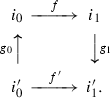(4.10)

Then for any $$\sigma ,\sigma ' \in {\text {Sec}}(I,\mathcal {C})$$ and $$f:i_0 \rightarrow i_1$$, let $${\mathcal {H}{} { om}}_f(\sigma ,\sigma ')$$ be the set of maps $$\widetilde{f}:\sigma (i_0) \rightarrow \sigma '(i_1)$$ such that $$\pi (\widetilde{f}) = f$$, and observe that a diagram (4.10) induces a map

\begin{aligned} {\text {Hom}}_f(\sigma ,\sigma ') \rightarrow {\text {Hom}}_{f'}(\sigma ,\sigma '), \qquad \widetilde{f} \mapsto \sigma '(g_1) \circ \widetilde{f} \circ \sigma (g_0), \end{aligned}

so that the sets $${\text {Hom}}_f(\sigma ,\sigma ')$$ patch together into a functor $${\mathcal {H}{} { om}}(\sigma ,\sigma ')$$ from $$\mathsf{A}(I)$$ to sets. We then have a natural identification

\begin{aligned} {\text {Hom}}(\sigma ,\sigma ') \cong \mathsf{lim}_{\mathsf{A}(I)}{\mathcal {H}{} { om}}(\sigma ,\sigma ') \end{aligned}
(4.11)

sending a map $$\varphi$$ to the collection of the elements $$f^*(\varphi (i')) \circ \sigma (f) = \sigma '(f) \circ \varphi (i) \in {\mathcal {H}{} { om}}_f(\sigma ,\sigma ')$$. If $$\pi$$ is constant, then this is the usual identification between homomorphisms and categorical “ends”, see e.g. [37, IX].

For any functor $$\gamma :I' \rightarrow I$$, the pullback $$\gamma ^*\mathcal {C}\rightarrow I'$$ is also a prefibration, with fibers $$(\gamma ^*\mathcal {C})_i = \mathcal {C}_{\gamma (i)}$$ and transition functors $$f^* = \gamma (f)^*$$. If I and $$I'$$ are small, then we have a natural pullback functor

\begin{aligned} \gamma ^*:{\text {Sec}}(I,\mathcal {C}) \rightarrow {\text {Sec}}(I',\gamma ^*\mathcal {C}). \end{aligned}
(4.12)

We will need two general results that allow one to construct an adjoint functor to (4.12)

### Lemma 4.16

Assume given a prefibration $$\mathcal {C}\rightarrow I$$ over a small category I, and another prefibration $$\gamma :I' \rightarrow I$$ from a small category $$I'$$. Moreover, assume that for any $$i \in I$$, limits $$\mathsf{lim}_{I'_i}$$ exist in the category $$\mathcal {C}_i$$, and for any morphism $$f:i \rightarrow i'$$, the transition functor $$f^*:\mathcal {C}_{i'} \rightarrow \mathcal {C}_i$$ commutes with these limits. Then the pullback functor (4.12) admits a right-adjoint functor

\begin{aligned} \gamma _*:{\text {Sec}}(I',\gamma ^*\mathcal {C}) \rightarrow {\text {Sec}}(I,\mathcal {C}), \end{aligned}
(4.13)

and for any $$i \in I$$, we have a natural isomorphism

\begin{aligned} \mathsf{ev}_i \circ \gamma _* \cong \mathsf{lim}_{I'_i} \circ e_i^*, \end{aligned}
(4.14)

where $$e_i:I'_i \rightarrow I'$$ is the embedding of the corresponding fiber of the prefibration $$\gamma$$.

### Proof

For any map $$f:i \rightarrow i'$$ in I and any section $$\sigma \in {\text {Sec}}(I',\gamma ^*\mathcal {C})$$, the maps (4.6) together provide a natural map

\begin{aligned} \left( f_\gamma ^*\right) ^*e_i^*\sigma \rightarrow f^*e_{i'}^*\sigma , \end{aligned}

where $$f_\gamma ^*:I'_{i'} \rightarrow I'_i$$ is the transition functor of the prefibration $$\gamma$$. Since $$f^*$$ commutes with limits, we then have a natural map

\begin{aligned} \mathsf{lim}_{I'_i}e_i^*\sigma \rightarrow \mathsf{lim}_{I'_{i'}}\left( f_\gamma ^*\right) ^*e_i^*\sigma \rightarrow \mathsf{lim}_{I'_{i'}}f^*e_{i'}^*\sigma \cong f^*\mathsf{lim}_{I'_{i'}}e_{i'}^*\sigma . \end{aligned}
(4.15)

Taking $$(\gamma _*\sigma )_i = \mathsf{lim}_{I'_i}e_i^*\sigma$$ with (4.15) as the maps (4.6) gives a well-defined section $$\gamma _*\sigma \in {\text {Sec}}(I,\mathcal {C})$$. The construction is obviously functorial in $$\sigma$$, this gives a functor (4.13) satisfying (4.14), and the adjunction maps $$\gamma ^* \circ \gamma _* \rightarrow \mathsf{Id}$$, $$\mathsf{Id}\rightarrow \gamma _* \circ \gamma ^*$$ are then induced by the adjunction maps for $$\mathsf{lim}_{I'_i}$$, $$i \in I$$. $$\square$$

For the second result, assume that we have two functors $$\gamma _0,\gamma _1:I' \rightarrow I$$ and a prefibration $$\mathcal {C}\rightarrow I$$. Observe that giving map $$a:\gamma _0 \rightarrow \gamma _1$$ is equivalent to giving a functor $$\widetilde{a}:I' \times  \rightarrow I$$ that restricts to $$\gamma _0$$ resp. $$\gamma _1$$ on $$I' \times 0 \subset I' \times $$ resp. $$I' \times 1 \subset I' \times $$.

### Definition 4.17

The map a is compatible with the prefibration $$\mathcal {C}$$ if for any morphism $$f:i \rightarrow i'$$ in $$I'$$, the natural map $$\gamma _0(f)^* \circ a(i')^* \rightarrow (a(i') \circ \gamma _0(f))^*$$ is an isomorphism, and strictly compatible with $$\mathcal {C}$$ if the maps $$a(i)^* \circ \gamma _1(f)^* \rightarrow (\gamma _1(f) \circ a(i))^*$$ are isomorphisms as well.

Then if a is compatible with $$\mathcal {C}$$, the projection $$\widetilde{a}^*\mathcal {C}\rightarrow $$ is a prefibration by Lemma 4.11, so that we are in the situation of Example 4.14. In particular, we have a natural functor

\begin{aligned} a^*:\gamma _1^*\mathcal {C}\rightarrow \gamma _0^*\mathcal {C}\end{aligned}
(4.16)

over $$I'$$ that evaluates to the transition functors $$a(i)^*$$ at every $$i \in I'$$. If a is strictly compatible with $$\mathcal {C}$$, then the functor (4.16) is cartesian over $$I'$$. If I and $$I'$$ are small, $$a^*$$ induces a functor on the categories of sections, and by the universal property of cartesian liftings, we have a natural morphism

\begin{aligned} \alpha (a):\gamma _0^* \rightarrow a^* \circ \gamma _1^* \end{aligned}
(4.17)

of functors from $${\text {Sec}}(I,\mathcal {C})$$ to $${\text {Sec}}(I',\gamma _0^*\mathcal {C})$$.

### Lemma 4.18

Assume given a functor $$\rho :I_0 \rightarrow I_1$$ between small categories, and a prefibration $$\pi :\mathcal {C}\rightarrow I_1$$. Moreover, assume that $$\rho$$ admits a left-adjoint functor $$\lambda :I_1 \rightarrow I_0$$, and the adjunction map $$a:\mathsf{Id}\rightarrow \rho \circ \lambda$$ is compatible with $$\mathcal {C}$$ in the sense of Definition 4.17. Then the pullback functor $$\rho ^*$$ of (4.12) admits a right-adjoint functor

\begin{aligned} \lambda ^\mathcal {C}:{\text {Sec}}(I_0,\rho ^*\mathcal {C}) \rightarrow {\text {Sec}}(I_1,\mathcal {C}) \end{aligned}

given by $$\lambda ^\mathcal {C}= a^* \circ \lambda ^*$$.

### Proof

By definition, for any prefibration $$\pi :\mathcal {C}\rightarrow I$$ over a small category I, we have a faithful embedding

\begin{aligned} \nu :{\text {Sec}}(I,\mathcal {C}) \hookrightarrow \mathcal {C}^I, \end{aligned}
(4.18)

and for any two sections $$\sigma ,\sigma ' \in {\text {Sec}}(I,\mathcal {C})$$, a map $$f:\nu (\sigma ) \rightarrow \nu (\sigma ')$$ comes from a map of sections if and only if $$\pi (f)=\mathsf{id}$$.

Consider now the categories $$\mathcal {C}^{I_0}$$, $$\mathcal {C}^{I_1}$$ of all functors from $$I_0$$ resp. $$I_1$$ to $$\mathcal {C}$$. Then the functors $$\lambda$$ and $$\rho$$ induce a pair of adjoint functors

\begin{aligned} \lambda ^*:\mathcal {C}^{I_0} \rightarrow \mathcal {C}^{I_1}, \qquad \rho ^*:\mathcal {C}^{I_1} \rightarrow \mathcal {C}^{I_0}, \end{aligned}

so that for any sections $$\sigma _0 \in {\text {Sec}}(I_0,\rho ^*\mathcal {C})$$, $$\sigma _1 \in {\text {Sec}}(I_1,\mathcal {C})$$, we have a natural isomorphism

\begin{aligned} {\text {Hom}}(\rho ^*\nu (\sigma _1),\nu (\sigma _0)) \cong {\text {Hom}}(\nu (\sigma _1),\lambda ^*\nu (\sigma _0)). \end{aligned}

Moreover, a map $$f:\rho ^*\nu (\sigma _1) \rightarrow \nu (\sigma _0)$$ comes from a map of sections if and only if $$\pi (f)=\mathsf{id}$$, and this is equivalent to saying that for the the adjoint map $$f_\dagger :\nu (\sigma _1) \rightarrow \lambda ^*\nu (\sigma _0)$$, we have $$\pi (f_\dagger )=a$$. It remains to observe that by by the universal property of the transition functors $$a(i)^*$$, $$i \in I_1$$, such maps are in one-to-on correspondence with maps from $$\sigma _1$$ to $$a^*\lambda ^*\sigma _0 = \lambda ^\mathcal {C}(\sigma _0)$$. $$\square$$

### Transpose fibrations

Let us now for the moment restrict our attention to fibrations and cofibrations. Then there is one additional general construction that will prove useful. Namely, observe that by definition, for any prefibration $$\mathcal {C}\rightarrow I$$, a map f in $$\mathcal {C}$$ can be factored as $$f = c \circ v$$ with cartesian c and vertical v, and such a factorization is unique up to a unique isomorphism. Moreover, for any cartesian $$c:c_1 \rightarrow c$$ and vertical $$v:c_2 \rightarrow c$$, there exists a cartesian square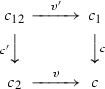(4.19)

in $$\mathcal {C}$$ with cartesian $$c'$$ and vertical $$v'$$. Dually, for a precofibration, we have cocartesian squares (4.19).

Now, if $$\mathcal {C}\rightarrow I$$ is a cofibration, define the transpose fibration $$\mathcal {C}^\perp \rightarrow I^o$$ as follows: its objects are objects of c, and morphisms from $$c_1$$ to $$c_2$$ are isomorphism classes of diagrams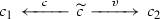(4.20)

in $$\mathcal {C}$$, with compositions obtained by taking the cocartesian squares (4.19). The fibers and the transition functors for $$\mathcal {C}^\perp \rightarrow I^o$$ are then exactly the same as for $$\mathcal {C}\rightarrow I$$, and the natural isomorphisms (4.2) are inverse to the corresponding isomorphisms (4.4) for $$\mathcal {C}\rightarrow I$$.

Analogously, for any fibration $$\mathcal {C}\rightarrow I$$, we can define the transpose cofibration $$\mathcal {C}_\perp \rightarrow I^o$$ by taking $$\mathcal {C}_\perp = (\mathcal {C}^{o\perp })^o$$. We then have natural equivalences

\begin{aligned} (\mathcal {C}^\perp )_\perp \cong \mathcal {C}, \qquad (\mathcal {C}_\perp )^\perp \cong \mathcal {C}. \end{aligned}
(4.21)

For a bifibration $$\mathcal {C}\rightarrow I$$, both $$\mathcal {C}_\perp$$ and $$\mathcal {C}^\perp$$ are well-defined and equipped with functors to $$I^o$$. For example, say that a fibration $$\mathcal {C}\rightarrow I$$ is locally constant if all its transition functors $$f^*$$ are equivalences. Then in particular, the functors $$f^*$$ have left-adjoint functors $$f_!$$, so that $$\mathcal {C}\rightarrow I$$ is a bifibration, and in this case, $$\mathcal {C}^\perp \cong \mathcal {C}_\perp \rightarrow I$$ is also a bifibration. For a general bifibration, $$\mathcal {C}_\perp$$ and $$\mathcal {C}^\perp$$ are completely different.

The transpose fibration is obviously functorial with respect to cocartesian functors, in that a cocartesian functor $$F:\mathcal {C}_1 \rightarrow \mathcal {C}_2$$ between two cofibrations $$\mathcal {C}_1,\mathcal {C}_2/I$$ indudes a cartesian functor $$F^\perp :\mathcal {C}_1^\perp \rightarrow \mathcal {C}_2^\perp$$, and dually for fibrations. In particular, for any fibration $$\mathcal {C}\rightarrow I$$ over a small category I, we have a natural equivalence

\begin{aligned} {\text {Sec}}_\natural (I,\mathcal {C}) \cong {\text {Sec}}^\natural (I^o,\mathcal {C}_\perp ), \end{aligned}
(4.22)

where $${\text {Sec}}_\natural$$, $${\text {Sec}}^\natural$$ are as in (4.8), (4.9), and dually for cofibrations.

Transpose fibrations arise naturally when one studies adjoints to functors over I. Namely, assume given two prefibrations $$\mathcal {C},\mathcal {C}' \rightarrow I$$, and a cartesian functor $$F:\mathcal {C}\rightarrow \mathcal {C}'$$ such that for any $$i \in I$$, F(i) admits a left-adjoint functor $$F(i)_\dagger$$. Then the functor F also has a left-adjoint functor

\begin{aligned} F_\dagger :\mathcal {C}' \rightarrow \mathcal {C}\end{aligned}
(4.23)

over I, with $$F_\dagger (i) = F(i)_\dagger$$, $$i \in I$$, and the maps (4.5) adjoint to the inverses of the isomorphisms (4.5) for the functor F. Dually, for any functor $$F:\mathcal {C}\rightarrow \mathcal {C}'$$ over I, assume that for any $$i \in I$$, F(i) has a right-adjoint functor $$F(i)^\dagger$$. Then for any morphism $$f:i \rightarrow i'$$, the map (4.5) induces by adjunction a natural map

\begin{aligned} f^* \circ F(i')^\dagger \rightarrow F(i)^\dagger \circ f^*, \end{aligned}
(4.24)

and if these maps are invertible, the inverse maps turn the collection $$F(i)^\dagger$$, $$i \in I$$ into a functor

\begin{aligned} F^\dagger :\mathcal {C}' \rightarrow \mathcal {C}\end{aligned}
(4.25)

over I right-adjoint to F. If the maps (4.24) are not invertible, the construction breaks down. However, assume that $$\mathcal {C},\mathcal {C}' \rightarrow I$$ are actually fibrations. Then what the maps (4.24) define is a functor

\begin{aligned} F^\dagger _\perp :\mathcal {C}_\perp \rightarrow \mathcal {C}'_\perp \end{aligned}
(4.26)

over $$I^o$$. If the maps (4.24) are isomorphisms, then $$F^\dagger _\perp = (F^\dagger )_\perp$$, where $$F^\dagger$$ is the adjoint functor of (4.25). In the general case, all one can say is that if I is small, so that we have the categories of sections and the equivalences (4.22), then for any $$\sigma \in {\text {Sec}}_\natural (I,\mathcal {C}) \cong {\text {Sec}}^\natural (I^o,\mathcal {C}_\perp )$$ and $$\sigma ' \in {\text {Sec}}_\natural (I,\mathcal {C}') \cong {\text {Sec}}^\natural (I^o,\mathcal {C}'_\perp )$$, we have a natural identification

\begin{aligned} {\text {Hom}}(\sigma ,F(\sigma ')) \cong {\text {Hom}}\left( F^\dagger _\perp (\sigma ),\sigma '\right) \end{aligned}
(4.27)

induced by the adjunctions between F(i) and $$F(i)^\dagger$$, $$i \in I$$.

All the statements and constructions on transpose fibrations and cofibrations are functorial with respect to I—that is, if we have a fibration resp. cofibration $$\mathcal {C}\rightarrow I$$ and a functor $$\gamma :I' \rightarrow I$$, then $$(\gamma ^*\mathcal {C})^\perp \cong \gamma ^*\mathcal {C}^\perp$$ resp. $$(\gamma ^*\mathcal {C})_\perp \cong \gamma ^*\mathcal {C}_\perp$$, and this is compatible with (4.21), (4.22), (4.26) and (4.27). In particular, assume that we have a commutative diagram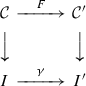(4.28)

of categories and functors such that the vertical row are fibrations, I and $$I'$$ are small, and for any $$i \in I$$, the functor $$F(i):\mathcal {C}_i \rightarrow \mathcal {C}'_{\gamma (i)}$$ has a right-adjoint $$F(i)^\dagger$$. Then we can factor F as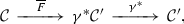and we can define a functor $$F^\perp :{\text {Sec}}(I^{'o},\mathcal {C}'_\perp ) \rightarrow {\text {Sec}}(I^o,\mathcal {C}_\perp )$$ as the composition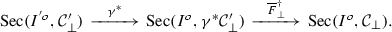This is compatible with compositions, in that we have a natural isomorphism $$(F_1 \circ F_2)^\perp \cong F_2^\perp \circ F_1^\perp$$ for a composable pair of diagrams (4.28).

This has one useful corollary. Assume that we have a cofibration with small fibers $$\gamma :I' \rightarrow I$$ and a prefibration $$\pi :\mathcal {C}\rightarrow I$$ that satisfy the assumptions of Lemma 4.12, so that $$\gamma \circ \pi :\mathcal {C}\rightarrow I$$ is also a cofibration and $$\pi$$ is cartesian over I. Then in general, the categories of sections $${\text {Sec}}(I_i,\mathcal {C}_i)$$ for different objects $$i \in I$$ are not related in any meaningful way. However, assume that for any $$i \in I$$, the prefibration $$\pi :\mathcal {C}_i \rightarrow I_i$$ is actually a fibration, with the transpose cofibration $$(\mathcal {C}_i)_\perp$$. Then for any morphism $$f:i \rightarrow i'$$ in I, the transition functors $$f^I_!$$, $$f^\mathcal {C}_!$$ of the cofibrations $$\gamma$$, $$\gamma \circ \pi$$ fit into a diagram (4.28), so that we obtain a natural functor

\begin{aligned} f^* = \left( f^\mathcal {C}_!\right) ^\perp :{\text {Sec}}\left( I^o_{i'},(\mathcal {C}_{i'})_\perp \right) \rightarrow {\text {Sec}}\left( I^o_i,(\mathcal {C}_i)_\perp \right) \end{aligned}

and isomorphisms $$(f_1 \circ f_2)^* \cong f_2^* \circ f_1^*$$ for a composable pairs of maps $$f_1$$, $$f_2$$. By the Grothedieck construction, this produces a fibration

\begin{aligned} \gamma ^\perp \mathcal {C}\rightarrow I \end{aligned}
(4.29)

with fibers $$(\gamma ^\perp \mathcal {C})_i \cong {\text {Sec}}(I^o_i,(\mathcal {C}_i)_\perp )$$. Moreover, assume that we have another prefibration $$\pi ':\mathcal {C}' \rightarrow I'$$ with the same properties, and a functor $$F:\mathcal {C}\rightarrow \mathcal {C}'$$ over $$I'$$ cocartesian over I such for for any $$i \in I'$$, the functor F(i) has a right-adjoint $$F(i)^\dagger$$. Then for any $$i \in I$$, F restricts to a functor $$F(i):\mathcal {C}_i \rightarrow \mathcal {C}'_i$$ over $$I_i$$, and the corresponding functors (4.26) together provide a functor

\begin{aligned} F^\perp :\gamma ^\perp \mathcal {C}' \rightarrow \gamma ^\perp \mathcal {C}\end{aligned}
(4.30)

cartesian over I.

### Categorical gluing

Extrapolating from Example 4.13, one could expect that for a general normalized covariant lax functor from some small category I to $${\text {Cat}}$$, the appropriate gluing is given by the category of sections $${\text {Sec}}(I,\mathcal {C})$$ of the corresponding precofibration $$\mathcal {C}\rightarrow I$$. However, this is not what happens. As a sign of this, we observe that Example 4.13 cannot be iterated: the maps (4.1) and (4.2) go in the opposite directions. Thus a collection of categories $$\mathcal {C}_i$$, $$i=0,1,2$$ and functors $$\Phi _{ij}:\mathcal {C}_i \rightarrow C_j$$, $$0 \le i <j \le 2$$ related by a map (4.1) form a precofibration over the category $$ = \{0,1,2\}$$, not a prefibration, and the category of sections of this precobiration has nothing to do with the iterated comma-category $$\mathsf{R}(\Phi _\cdot )$$.

To find the correct notion of a good gluing, it is convenient to axiomatize the situation. Assume given a category I. Denote by $$I^<$$ the category obtained by formally adding an initial object o to I, and denote by $$j:I \rightarrow I^<$$ the natural embedding functor.

### Definition 4.19

A 2-limit diagram over I is a precofibration $$\mathcal {C}^< \rightarrow I^<$$ such that for any precofibration $$\widetilde{\mathcal {C}} \rightarrow I^<$$, any equivalence $$\rho :j^*\widetilde{\mathcal {C}} \rightarrow j^*\mathcal {C}^<$$ over $$I \subset I^<$$ extends to a functor $$\widetilde{\rho }:\widetilde{\mathcal {C}} \rightarrow \mathcal {C}^<$$ over $$I^<$$ cocartesian over maps $$o \rightarrow i$$, $$i \in I$$, and this extension is unique up to a unique isomorphism.

### Lemma 4.20

For any precofibration $$\mathcal {C}\rightarrow I$$ over a small category I, there exists a 2-limit diagram $$\mathcal {C}^< \rightarrow I^<$$ equipped with an equivalence $$j^*\mathcal {C}^< \cong \mathcal {C}$$ over I. Moreover, any two such 2-limit diagrams are equivalent over $$I^<$$, and the equivalence is unique up to a unique isomorphism.

### Proof

The uniqueness claims follow immediately from the universal property of Definition 4.19. To prove existence, choose a cleavage of the precofibration $$\mathcal {C}\rightarrow I$$, thus fixing the transition functors $$f_!$$ and the maps (4.4). For any object $$i \in I$$, denote by $$o(i):o \rightarrow i$$ the unique map. To construct $$\mathcal {C}^<$$, we need to construct the fiber $$\mathcal {C}^<_o$$ over the initial object $$o \in I^<$$, a functor $$o(i)_!:\mathcal {C}^<_o \rightarrow \mathcal {C}_i$$ for any object $$i \in I$$, and a morphism $$\alpha (f,o(i)):o(i')_! \rightarrow f_! \circ o(i)_!$$ for any morphism $$f:i \rightarrow i'$$ in I, such that $$\alpha (o(i),\mathsf{id})=\mathsf{id}$$, and the squares (4.3) are commutative (that is, $$(\alpha (f,f') \circ \mathsf{id}) \circ \alpha (o(i),f' \circ f) = (\mathsf{id}\circ \alpha (o(i),f)) \circ \alpha (f',o(i'))$$ for any morphisms $$f:i \rightarrow i'$$, $$f':i' \rightarrow i''$$ in I). We let $$\mathcal {C}^<_o$$ be the category of the following data:

1. (i)

an object $$\rho (i) \in \mathcal {C}_i$$ for any object $$i \in I$$, and

2. (ii)

a morphism $$\rho (f):\rho (i') \rightarrow f_!\rho (i)$$ for any morphism $$f:i \rightarrow i'$$ in I,

such that $$\rho (\mathsf{id})=\mathsf{id}$$ and $$\rho (f) \circ \rho (f') = \alpha (f',f) \circ \rho (f' \circ f)$$ for any morphisms $$f:i \rightarrow i'$$, $$f':i' \rightarrow i''$$ in I). The functor $$o(i)_!$$ then sends a collection $$\langle \rho _\cdot \rangle$$ to $$\rho (i)$$, and the morphism $$\alpha (f,o(i))$$ is the morphism $$\rho (f)$$. $$\square$$

### Definition 4.21

For any precofibration $$\pi :\mathcal {C}\rightarrow I$$ over a small category I with the 2-limit diagram $$\mathcal {C}^< \rightarrow I^<$$ provided by Lemma 4.20, the fiber $$\mathcal {C}^<_o$$ over the initial object $$o \in I^<$$ is denoted $${\text {Rec}}(I,\mathcal {C})$$, where $${\text {Rec}}$$ stands for “recollement”, and its objects are called cosections of the precofibration $$\pi$$.

Explicitly, cosections are described by the data (i), (ii) in the proof of Lemma 4.20. Note that by definition, for any precofibration $$\mathcal {C}\rightarrow I^<$$, we have a natural functor $$\Phi :\mathcal {C}_o \rightarrow {\text {Rec}}(I,j^*\mathcal {C})$$, and it immediately follows from the explicit description that we have a natural equivalence

\begin{aligned} {\text {Rec}}(I^<,\mathcal {C}) \cong \mathsf{R}(\Phi ), \end{aligned}
(4.31)

where $$\mathsf{R}(\Phi )$$ is the right-comma category for the functor $$\Phi$$. In particular, for any $$n \ge 0$$ we have $$[n]^< \cong [n+1]$$, so that for any precofibration $$\mathcal {C}\rightarrow [n]$$, $${\text {Rec}}([n],\mathcal {C})$$ is indeed obtained by iterating the comma-category construction.

If the precofibration $$\mathcal {C}\rightarrow I$$ is in fact a cofibration, with the transpose fibration $$\mathcal {C}^\perp \rightarrow I$$, then we have a natural equivalence of categories

\begin{aligned} {\text {Rec}}(I,\mathcal {C}) \cong {\text {Sec}}(I^o,\mathcal {C}^\perp ) \end{aligned}
(4.32)

that sends a cosection $$\langle \rho _\cdot \rangle$$ to the section given by $$\sigma (i)=\rho (i)$$, $$\sigma (f) = \rho (f)$$. However, in the general case, the only description of cosections of $$\mathcal {C}\rightarrow I$$ that we have at the moment is in terms of the data (i), (ii) in the proof of Lemma 4.20.

We note that this explicit can be easily modified to apply to an arbitrary covariant lax functor, nor only to the normalized ones. The resulting category of cosections would not satisfy any obvious universal property, but it will be well-defined. We will not do it in the general case. Instead, let us consider another very well-known special case—namely, the case when I is the point category $$\mathsf{pt}$$. Covariant lax functors from $$\mathsf{pt}$$ to $${\text {Cat}}$$ are known as comonads.

Recall that explicitly, a comonad on a category $$\mathcal {C}$$ is a functor $$\Phi :\mathcal {C}\rightarrow \mathcal {C}$$ equipped with two maps $$\varepsilon :\Phi \rightarrow \mathsf{Id}$$, $$\mu :\Phi \rightarrow \Phi \circ \Phi$$ such that $$(\varepsilon \circ \mathsf{id}) \circ \mu = (\mathsf{id}\circ \varepsilon ) \circ \mu = \mathsf{id}$$ and $$(\mu \circ \mathsf{id}) \circ \mu = (\mathsf{id}\circ \mu ) \circ \mu$$ (if $$\mathcal {C}$$ is small, one can equivalently say that $$\Phi$$ is a coalgebra in the monoidal category of endofunctors of $$\mathcal {C}$$). A standard source of comonads is adjunction: a functor $$\rho :\mathcal {C}\rightarrow \mathcal {E}$$ to some category $$\mathcal {C}$$ that admits a left-adjoint $$\lambda :\mathcal {E}\rightarrow \mathcal {C}$$ induces a comonad structure on $$\Phi = \lambda \circ \rho$$. Dually, $$\Phi _\dagger = \rho \circ \lambda$$ is a monad on $$\mathcal {E}$$ (that is, a comonad on the opposite category $$\mathcal {E}^o$$).

A coalgebra over a comonad $$\Phi$$ is an object $$X \in \mathcal {C}$$ equipped with a map $$\alpha :X \rightarrow \Phi (X)$$ such that $$\varepsilon \circ \alpha = \mathsf{id}$$ and $$\Phi (\alpha ) \circ \alpha = \mu \circ \alpha$$. Coalgebras over $$\Phi$$ form a category, denoted $${\text {Coalg}}(\mathcal {C},\Phi )$$, we have a forgetful functor $$\lambda :{\text {Coalg}}(\mathcal {C},\Phi ) \rightarrow \mathcal {C}$$, and it has a right-adjoint $$\rho :\mathcal {C}\rightarrow {\text {Coalg}}(\mathcal {C},\Phi )$$ sending $$X \in \mathcal {C}$$ to $$\Phi (X)$$ with the cofree coalgebra structure. The comonad induced by this adjoint pair is canonically identified with $$\Phi$$. The essential image $${\text {coalg}}(\mathcal {C},\Phi ) \subset {\text {Coalg}}(\mathcal {C},\Phi )$$ of the functor $$\rho$$ is known as the Kleisli category of the comonad; its objects are objects of $$\mathcal {C}$$, and we have

\begin{aligned} {\text {Hom}}_{{\text {coalg}}(\mathcal {C},\Phi )}(X,Y) = {\text {Hom}}_\mathcal {C}(\Phi (X),Y) \end{aligned}

for any $$X,Y \in \mathcal {C}$$. The categories $${\text {coalg}}(\mathcal {C},\Phi )$$ resp. $${\text {Coalg}}(\mathcal {C},\Phi )$$ are the initial resp. the terminal among all the categories generating the comonad $$\Phi$$ by adjunction: for any $$\mathcal {E}$$ with adjoint pair $$\rho :\mathcal {C}\rightarrow \mathcal {E}$$, $$\lambda :\mathcal {E}\rightarrow \mathcal {C}$$ generating the comonad $$\Phi$$, we have natural functors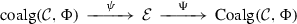(4.33)

that commute with $$\lambda$$ and $$\rho$$. The functor $$\Psi$$ sends $$e \in \mathcal {E}$$ to $$\lambda (e)$$ equipped with the map $$\lambda (a):\lambda (e) \rightarrow \Phi (\lambda (e)) = \lambda (\Phi _\dagger (e))$$, where $$a:e \rightarrow \Phi _\dagger (e)$$ is the adjunction map. Thus $${\text {Coalg}}(\mathcal {C},\Phi )$$ has a universal property similar to that of Definition 4.19, and can be thought of as a certain 2-limit in the category of categories.

### Example 4.22

Let $$\Delta$$ be the category of finite ordinals, as in Example 4.7 and Remark 4.8, and let $$\Delta _+ \subset \Delta$$ be the subcategory with the same objects and maps between them that send the initial element to the initial element. Then the embedding functor $$\rho :\Delta _+ \rightarrow \Delta$$ admits a left-adjoint $$\lambda :\Delta \rightarrow \Delta _+$$ that adds a new initial element to an ordinal. We thus obtain a comonad $$\varphi = \rho \circ \lambda$$ on $$\Delta _+$$. In this case, we have $${\text {coalg}}(\Delta _+,\varphi ) \cong \Delta$$, and $${\text {Coalg}}(\Delta _+,\varphi ) \supset {\text {coalg}}(\Delta _+,\varphi ) \cong \Delta$$ is the category $$\Delta ^<$$ obtained by adding the new initial element o corresponding to the empty ordinal $$\emptyset$$.

### Example 4.23

A real-life example of the comparison functor $$\Psi$$ of (4.33) is given by the Stein factorization in algebraic geometry. Namely, assume given a proper map $$f:X \rightarrow Y$$ of algebraic varieties, and consider the corresponding pair of adjoint functors $$f_*$$, $$f^*$$ between the categories of coherent sheaves $${\text {Coh}}(X)$$, $${\text {Coh}}(Y)$$. Then $$\Phi =f_* \circ f^*$$ is a monad on $${\text {Coh}}(Y)$$—that is, a comonad on the opposite category $${\text {Coh}}(Y)^o$$, We then have $${\text {Coalg}}({\text {Coh}}(Y)^o,\Phi ^o) \cong {\text {Coh}}(Y')^o$$, where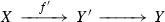is the Stein factorization of the map f, and we have $$\Psi \cong f'_*$$.

If we have a comonad $$\varphi$$ on a small category I, then for any category $$\mathcal {C}$$, $$\varphi ^*$$ is a comonad on the category of functors $$\mathcal {C}^I$$, and we have an obvious natural identification

\begin{aligned} {\text {Coalg}}(\mathcal {C}^I,\varphi ^*) \cong \mathcal {C}^{{\text {coalg}}(I,\varphi )}. \end{aligned}
(4.34)

We will need the following refinement of this identification.

### Lemma 4.24

In the assumptions of Lemma 4.18, denote by $$\varphi =\lambda \circ \rho$$ resp. $$\Phi = \lambda ^\mathcal {C}\circ \rho ^*$$ the comonads on $$I_0$$ resp. $${\text {Sec}}(I_0,\rho ^*\mathcal {C})$$ induced by the adjunction, and assume that the comparison functor $$\psi :{\text {coalg}}(I_0,\varphi ) \rightarrow I_1$$ of (4.33) is an equivalence. Then the comparison functor

\begin{aligned} \Psi :{\text {Sec}}(I_1,\mathcal {C}) \rightarrow {\text {Coalg}}({\text {Sec}}(I_0,\rho ^*\mathcal {C}),\Phi ) \end{aligned}

of (4.33) is also an equivalence.

### Proof

Let $$\varepsilon :\varphi \rightarrow \mathsf{id}$$, $$\mu :\varphi \rightarrow \varphi \circ \varphi$$ be the counit and comultiplication maps for the comonad $$\varphi$$, let $$\varphi _\dagger = \rho \circ \lambda$$ be the dual monad on $$I_1$$, and as in Lemma 4.18, let $$a:\mathsf{id}\rightarrow \varphi _\dagger$$ be the adjunction map. Note that we have

\begin{aligned} \begin{aligned} (\rho \circ \varepsilon ) \circ (a \circ \rho )&= \mathsf{id},\\ (\rho \circ \mu ) \circ (a \circ \rho )&= (a \circ \varphi \circ \rho ) \circ (a \circ \rho ), \end{aligned} \end{aligned}
(4.35)

and since a is assumed to be compatible with $$\mathcal {C}$$, we also have

\begin{aligned} ((a \circ \varphi \circ \rho ) \circ (a \circ \rho ))^* \cong (a \circ \rho )^* \circ (a \circ \varphi \circ \rho )^*. \end{aligned}
(4.36)

Consider now a section $$\sigma \in {\text {Sec}}(I_0,\rho ^*\mathcal {C})$$, and let $$\nu (\sigma ):I_0 \rightarrow \mathcal {C}$$ be its image under the embedding (4.18). Then by definition, a structure of a $$\Phi$$-coalgebra on $$\sigma$$ is given by a map $$\alpha :\sigma \rightarrow \Phi (\sigma )$$, and as in the proof of Lemma 4.18, such maps are in one-to-one correspondence with maps $$\widetilde{\alpha }:\nu (\sigma ) \rightarrow \nu (\sigma ) \circ \varphi$$ that project to the adjunction map $$a(\rho ):\rho \rightarrow \rho \circ \varphi$$ under the projection $$\pi :\mathcal {C}\rightarrow I_1$$. Moreover, such a map $$\widetilde{\alpha }$$ turns $$\nu (\sigma )$$ into a coalgebra over $$\varphi ^*$$ if and only if

\begin{aligned} \begin{aligned} \varepsilon \circ \widetilde{\alpha }&= \mathsf{id}:\nu (\sigma ) \rightarrow \nu (\sigma )),\\ \mu \circ \widetilde{\alpha }&= (\widetilde{\alpha } \circ \varphi ) \circ \widetilde{\alpha }:\nu (\sigma ) \rightarrow \nu (\sigma ) \circ \varphi \circ \varphi , \end{aligned} \end{aligned}

and by (4.35) and (4.36), this happens if and only if $$\alpha$$ turns $$\sigma$$ into a coalgebra over the comonad $$\Phi$$. By (4.34), $$\langle \nu (\sigma ),\widetilde{\alpha } \rangle$$ then defines a functor $$I_1 \rightarrow \mathcal {C}$$ that is a section of the prefibration $$\mathcal {C}$$. $$\square$$

## Simplicial replacements I

The categorical gluing constructions of Sect. 4.5 do not work too well in the homotopical setting: the associativity constraints involved in the definitions tend to produce higher order operations, and the whole assembly becomes very hard to control. Therefore we need another description of the categories $${\text {Rec}}(I,\mathcal {C})$$ and $${\text {Coalg}}(\mathcal {C},\Phi )$$. It turns out that this is possible if one uses simplicial methods, in the spirit of the treatment of monads and comonads in [37, VII.6]. In this section, we prepare the ground by introducing and studying a special class of prefibrations over the category $$\Delta$$ of Example 4.22.

### Special prefibrations

Say that a map $$f:[n] \rightarrow [n']$$ in the category $$\Delta$$ is special if $$f(0)=0$$ (that is, f is a map in the subcategory $$\Delta _+ \subset \Delta$$ of Example 4.22), and say that f is co-special if it is an isomorphism between [n] and a terminal segment of the ordinal $$[n']$$ (that is, $$f(i) = i+n'-n$$ for any $$i \in [n]$$). Observe that for any special map $$p:[n] \rightarrow [n_1]$$ and co-special map $$i:[n] \rightarrow [n_2]$$, we have a cocartesian square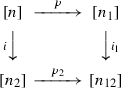(5.1)

with special $$p_2$$ and co-special $$i_1$$ (explicitly, $$n_{12} = n_1 + n_2 - n$$). We will call squares of this form standard. Moreover, every map $$f:[n] \rightarrow [n']$$ in $$\Delta$$ uniquely factors as

\begin{aligned} f = p \circ i \end{aligned}
(5.2)

with special i and co-special p, and there exists a unique standard square (5.1) with $$[n'] = [n_{12}]$$, $$p=p$$, $$i_1=i$$ (explicitly, $$[n_1]$$ is the terminal segment $$\{f(0),\dots ,n'\}$$ of the ordinal $$[n']$$, $$n_2 = n' + n - n_1$$, and $$p_2=\mathsf{id}$$ on $$\{0,\dots ,f(0)\} \subset [n_2]$$).

More generally, assume given a simplicial set $$X \in \Delta ^o{\text {Sets}}$$, and denote by $$\Delta X$$ its category of simplices—its objects are pairs $$\langle [n],x \rangle$$, $$[n] \in \Delta$$, $$x \in X([n])$$, and morphisms from $$\langle [n],x \rangle$$ to $$\langle [n'],x' \rangle$$ are morphisms $$f:[n] \rightarrow [n']$$ such that $$f(x')=x$$. The forgetful functor $$\pi :\Delta X \rightarrow \Delta$$, $$\langle [n],x \rangle \mapsto [n]$$ is the discrete fibration that corresponds to X by the Grothendieck construction, and every discrete fibration over $$\Delta$$ with small fibers arises in this way.

Since $$\pi :\Delta X \rightarrow \Delta$$ is a discrete fibration, lifting a morphism $$f:[n] \rightarrow [n']$$ in $$\Delta$$ to a morphism in $$\Delta X$$ amounts to choosing a simplex $$x' \in X([n'])$$, and lifting a commutative square (5.1) to a commutative square in $$\Delta X$$ amounts to choosing $$x_{12} \in X([n_{12}])$$. Say that such a lifted morphism f is special resp. co-special if it is so as a morphism in $$\Delta$$, and say such a lifted square is standard if it is standard in $$\Delta$$.

### Definition 5.1

For any $$X \in \Delta ^o{\text {Sets}}$$, a prefibration $$\mathcal {C}\rightarrow \Delta X$$ is special if

1. (i)

for any special map f in $$\Delta$$, $$f^*$$ is an equivalence of categories,

2. (ii)

the natural map (4.2) is an isomorphism as soon as both $$f_1$$ and $$f_2$$ are either special or co-special, and

3. (iii)

for any standard square (5.1) with $$f=i_1 \circ p = i \circ p_1$$, the maps (4.2) provide isomorphisms $$p^* \circ i_1^* \cong f^* \cong i^* \circ p_1^*$$.

A special prefibration $$\pi :\mathcal {C}\rightarrow \Delta X$$ is normalized if in addition,

1. (iv)

the natural map (4.2) is an isomorphism if $$\pi (f_1)$$ is surjective.

A section of such a prefibration is special if it is cartesian along all special maps in the sense of Definition 4.5 (ii), and $${\text {Sec}}_+(\Delta X,\mathcal {C}) \subset {\text {Sec}}(\Delta X,\mathcal {C})$$ is the full subcategory spanned by special sections.

### Remark 5.2

Note that since $$ \in \Delta _+ \subset \Delta$$ is the initial object of the category $$\Delta _+$$, for any simplicial set X and object $$c \in \Delta X$$, there exists a unique special map $$s:c' \rightarrow c$$ such that $$\pi (c') =  \in \Delta$$. We will call such maps base special maps. Then Definition 5.1 (i),(ii) immediately show that it suffices to check Definition 5.1 (iii) for squares (5.1) such that p is a base special map.

For an example of a special fibration, assume given a small category I, and denote by $$\Delta I = \Delta NI$$ the category of simplices of its nerve NI ($$\Delta I$$ is also known as the simplicial replacement of I, see e.g.  or ). Explicitly, objects in $$\Delta I$$ are pairs $$\langle [n],i_\cdot \rangle$$ of an object $$[n] \in \Delta$$ and a diagram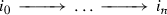(5.3)

in I. Sending a pair $$\langle [n],i_\cdot \rangle$$ to $$i_0$$ defines a functor

\begin{aligned} \xi :\Delta I \rightarrow I^o \end{aligned}
(5.4)

that inverts all special maps in $$\Delta I$$. Then for any cofibration $$\mathcal {C}\rightarrow I$$ with the transpose fibration $$\mathcal {C}^\perp$$, the pullback $$\xi ^*\mathcal {C}^\perp$$ is a special fibration over $$\Delta I$$ in the sense of Definition 5.1, and we have a pullback functor

\begin{aligned} \xi ^*:{\text {Rec}}(I,\mathcal {C}) \cong {\text {Sec}}(I,\mathcal {C}^\perp ) \rightarrow {\text {Sec}}_+(\Delta I,\xi ^*\mathcal {C}^\perp ). \end{aligned}
(5.5)

Constructing special prefibrations is more difficult (in particular, the pullback $$\xi ^*\mathcal {C}'$$ of a prefibration $$\mathcal {C}' \rightarrow I^o$$ in general is not special). Here is one simple result that helps.

### Definition 5.3

For any simplicial set X, a fibration $$\mathcal {C}' \rightarrow \Delta X$$ is called generating if

1. (i)

for any base special map $$f:c' \rightarrow c$$ in $$\Delta X$$, the corresponding transition functor $$f^*:\mathcal {C}'_c \rightarrow \mathcal {C}'_{c'}$$ admits a fully faithful left-adjoint $$f_!:\mathcal {C}'_{c'} \rightarrow \mathcal {C}'_c$$ with essential image $$\mathcal {C}_c \subset \mathcal {C}'_c$$, and

2. (ii)

for any co-special map $$f:c \rightarrow c'$$ in $$\Delta X$$, the corresponding transition functor $$f^*:\mathcal {C}'_{c'} \rightarrow \mathcal {C}'_c$$ sends $$\mathcal {C}_{c'} \subset \mathcal {C}'_{c'}$$ into $$\mathcal {C}_c \subset \mathcal {C}'_c$$.

### Lemma 5.4

Assume given a simplicial set X and a generating fibration $$\mathcal {C}' \rightarrow \Delta X$$, and let $$\mathcal {C}\subset \mathcal {C}'$$ be the full subcategory spanned by the subcategories $$\mathcal {C}_c \subset \mathcal {C}'_c \subset \mathcal {C}'$$, $$c \in \Delta X$$. Then the projection $$\mathcal {C}\rightarrow \Delta$$ is a special prefibration in the sense of Definition 5.1, and the embedding $$\mathcal {C}\rightarrow \mathcal {C}'$$ is cartesian along co-special and base special maps

### Proof

The fact that $$\mathcal {C}\rightarrow \Delta$$ is a prefibration and $$\mathcal {C}\rightarrow \mathcal {C}'$$ is cartesian along base special maps immediately follows from Definition 5.3 (i), as in Example 4.9. Moreover, since $$f_!$$ is fully faithful, the transition functor $$f^*:\mathcal {C}_c \rightarrow \mathcal {C}_{c'}$$ is an equivalence for any base special map $$f:c' \rightarrow c$$, and for any special map $$g:c \rightarrow c''$$, we have $$g^* \circ f_! \cong (g \circ f)_!$$. Thus $$(g \circ f)^* \cong f^* \circ g^*$$, so that $$f^*$$ is an equivalence, and we have $$(f_1 \circ f_2)^* \cong f_2^* \circ f_1^*$$ for any composable pair of special maps $$f_1$$, $$f_2$$. This proves Definition 5.1 (i) and the special case of (ii). Then by Definition 5.3 (ii), for any co-special map $$f:c \rightarrow c'$$, the transition functor $$f^*:\mathcal {C}'_{c'} \rightarrow \mathcal {C}'_c$$ of the fibration $$\mathcal {C}'$$ restricts to the transition functor of the prefibration $$\mathcal {C}$$. This means that $$\mathcal {C}\rightarrow \mathcal {C}'$$ is cartesian along f. Moreover, since $$\mathcal {C}'$$ is a fibration, this implies the remaining co-special case of Definition 5.1 (ii), and Definition 5.1 (iii) for a square (5.1) with base special map p. By Remark 5.2, this is enough. $$\square$$

### Canonical embedding

It turns out that Lemma 5.4 admits a converse: every special prefibration $$\mathcal {C}\rightarrow \Delta X$$ is canonically generated by a generating fibration $$\mathcal {C}' \rightarrow \Delta X$$. To prove this, we need one useful generalization of the simplicial replacement construction.

Say that a class of morphisms v in a category $$\mathcal {C}$$ is multiplicative if it is closed under compositions and contains all identity maps, and assume given a category $$\mathcal {C}$$ and two classes of morphisms v, c in $$\mathcal {C}$$ such that v is multiplicative.

### Definition 5.5

The simplicial expansion $$\Delta ^c_v \mathcal {C}$$ of the category $$\mathcal {C}$$ with respect to the classes v, c is the category such that

• objects of $$\Delta ^c_v \mathcal {C}$$ are pairs $$\langle [n],\kappa \rangle$$ of an ordinal $$[n] \in \Delta$$ and a functor $$\kappa :[n] \rightarrow \mathcal {C}$$ such that for any $$l \in [n]$$, $$l \ge 1$$, the map $$\kappa (l-1) \rightarrow \kappa (l)$$ is in the class c, and

• morphisms from $$\langle [n],\kappa \rangle$$ to $$\langle [n'],\kappa ' \rangle$$ are pairs $$\langle f,b \rangle$$ of a map $$f:[n] \rightarrow [n']$$ in $$\Delta$$ and a map $$b:\kappa \rightarrow \kappa ' \circ f$$ such that for any $$i \in [n]$$, the map $$b(i):\kappa (i) \rightarrow \kappa '(f(i))$$ is in the class v.

Note that for any map $$\langle f,b \rangle :\langle [n],\kappa \rangle \rightarrow \langle [n'],\kappa ' \rangle$$ in $$\Delta ^c_v\mathcal {C}$$, we have a natural composition map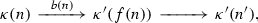(5.6)

and sending $$\langle [n],\kappa \rangle$$ to $$\kappa (n)$$ and $$\langle f,b \rangle$$ to the map (5.6) then gives a natural functor

\begin{aligned} \beta :\Delta ^c_v\mathcal {C}\rightarrow \mathcal {C}. \end{aligned}
(5.7)

If c is the class $$\tau$$ of all maps in $$\mathcal {C}$$, or if v is the class $$\mathsf{id}$$ of identity maps, we will drop it from notation, so that $$\Delta _v\mathcal {C}= \Delta ^\tau _v\mathcal {C}$$ and $$\Delta \mathcal {C}=\Delta ^\tau _{\mathsf{id}}\mathcal {C}$$ (in particular, $$\Delta I$$ for a small category I is its simplicial replacement, so that our notation is consistent). For any c, v, we have a natural forgetful functor $$\Delta ^v_c \mathcal {C}\rightarrow \Delta$$. If c is multiplicative, this functor is a fibration, with transition functor $$f^*$$ for a map $$f:[n'] \rightarrow [n]$$ sending $$\langle [n],\kappa \rangle$$ to $$\langle [n'], \kappa \circ f \rangle$$.

Now assume given a simplicial set X and a special prefibration $$\pi :\mathcal {C}\rightarrow \Delta X$$. Say that a map f in $$\mathcal {C}$$ is special if $$\pi (f)$$ is special, and say that f is co-special if $$\pi (f)$$ is co-special and f is cartesian over $$\Delta$$. Denote the classes of special resp. co-special maps by s resp. c. Note that the class s is multiplicative, and by Definition 5.1 (ii), the same is true for the class c. Denote by $$\mathcal {C}_+,\mathcal {C}_- \subset \mathcal {C}$$ the subcategories spanned by special resp. co-special maps.

### Lemma 5.6

For any special prefibration $$\mathcal {C}\rightarrow \Delta X$$, the functor $$\beta :\Delta ^c_s \mathcal {C}\rightarrow \mathcal {C}$$ of (5.7) admits a fully faithful right-adjoint functor

\begin{aligned} A:\mathcal {C}\rightarrow \Delta ^c_s\mathcal {C}. \end{aligned}
(5.8)

### Proof

Definition 5.1 (iii) immediately implies that any map f in $$\mathcal {C}$$ has a decomposition (5.2) with special i and co-special p. Therefore for any object $$c \in \mathcal {C}$$ and any object $$\langle [n],\kappa \rangle \in \widetilde{\mathcal {C}}$$, a map $$\kappa (n) \rightarrow c$$ induces a map $$\kappa (i) \rightarrow c$$ for any $$i \in [n]$$, and it decomposes as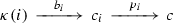with special $$b_i$$ and co-special $$p_i$$. Then sending $$i \in [n]$$ to $$p_i:c_i \rightarrow c$$ gives a functor $$f:[n] \rightarrow \mathcal {C}_-/c$$, where $$\mathcal {C}_-/c$$ is category of objects $$c' \in \mathcal {C}$$ equipped with a co-special map $$c' \rightarrow c$$, and the maps $$b_i$$ define a pointwise-special map $$b:\kappa \rightarrow \tau (c)$$, where $$\tau (c):\mathcal {C}_-/c \rightarrow c$$ sends $$c' \rightarrow c$$ to $$c'$$. To construct the adjoint functor (5.8), it suffices to observe that the $$\mathcal {C}_-/c$$ is naturally equivalent to $$\pi (c) \in \Delta$$, and let $$A(c) = \langle \pi (c),\tau (c) \rangle$$. We also see that $$\beta \circ A \cong \mathsf{id}$$, so that A is fully faithful. $$\square$$

Consider now the category $$\Delta _\flat$$ of pairs $$\langle [n],l \rangle$$ described in Example 4.7, with its cofibration $$\nu :\Delta _\flat \rightarrow \Delta$$, $$\langle [n],l \rangle \mapsto [n]$$. Then $$\nu$$ admits a fully faithful right-adjoint functor $$\sigma :\Delta \rightarrow \Delta _\flat$$, $$[n] \mapsto \langle [n],0 \rangle$$, and it in turn has a right-adjoint functor $$\sigma _\dagger :\Delta _\flat \rightarrow \Delta$$ that sends $$\langle [n],l \rangle$$ to the ordinal $$\{l,\dots ,n\rangle$$. Moreover, for any simplicial set X, let $$\Delta _\flat X = \Delta _\flat \times _\Delta \Delta X$$, with the induced cofibration $$\nu :\Delta _\flat X \rightarrow \Delta X$$. Then $$\nu$$ still has a fully faithful right-adjoint functor $$\sigma$$ sending $$\langle [n],x \rangle$$ to $$\langle [n],0,x \rangle$$, and it has a right-adjoint functor $$\sigma _\dagger$$ given by

\begin{aligned} \sigma _\dagger (\langle [n],l,x \rangle ) = \langle \sigma _\dagger (\langle [n],l\rangle ),a^*x \rangle , \end{aligned}
(5.9)

where $$a:\sigma (\sigma _\dagger (\langle [n],l \rangle )) \rightarrow \langle [n],l \rangle$$ is the adjunction map. Furthermore, for any map f in $$\Delta _\flat X$$ that is vertical resp. cocartesian with respect to $$\nu$$, the map $$\sigma _\dagger (f)$$ in $$\Delta X$$ is co-special resp. special. Therefore by Definition 5.1 (i),(iii), for any special prefibration $$\mathcal {C}\rightarrow \Delta X$$, the induced prefibration $$\sigma _\dagger ^*\mathcal {C}\rightarrow \Delta _\flat X$$ and the cofibration $$\nu :\Delta _\flat X \rightarrow \Delta X$$ satisfy the assumptions of Lemma 4.12. Moreover, by Definition 5.1 (ii), $$\sigma _\dagger ^*\mathcal {C}$$ restricts to a fibration on every fiber of $$\nu :\Delta _\flat X \rightarrow \Delta X$$, so that we have a well-defined fibration

\begin{aligned} B(\mathcal {C}) \cong \nu ^\perp \sigma _\dagger ^*\mathcal {C}\rightarrow \Delta X \end{aligned}
(5.10)

provided by (4.30).

### Lemma 5.7

The fibration $$B(\mathcal {C}) \rightarrow \Delta X$$ of (5.10) is generating in the sense of Definition 5.3, and the corresponding special prefibration over $$\Delta X$$ is naturally equivalent to $$\mathcal {C}$$.

### Proof

By definition, the fiber $$B(\mathcal {C})_{\langle [n],x \rangle }$$ of the fibration (5.10) over an object $$\langle [n],x \rangle \in \Delta X$$ is given by

\begin{aligned} B(\mathcal {C})_{\langle [n],x \rangle } \cong {\text {Sec}}([n],(\sigma _n^*\mathcal {C})_\perp ), \end{aligned}
(5.11)

where $$\sigma _n:[n]^o \rightarrow \Delta X$$ is the restriction of the functor $$\sigma _\dagger$$ to the fiber $$[n]^o \cong \nu ^{-1}(\langle [n],x \rangle ) \subset \Delta _\flat X$$. Moreover, the transition functor $$s^*$$ with respect to a base special map $$s:\langle ,s^*x \rangle \rightarrow \langle [n],x \rangle$$ is given by evaluation at the initial object $$0 \in [n]$$. For any cofibration $$\mathcal {E}\rightarrow [n]$$, evaluation at 0 has a fully faithful left-adjoint functor $$\mathcal {E}_0 \rightarrow {\text {Sec}}([n],\mathcal {E})$$ whose image is the subcategory $${\text {Sec}}^\natural ([n],\mathcal {E}) \subset {\text {Sec}}([n],\mathcal {E})$$ of cocartesian sections. Therefore $$B(\mathcal {C})$$ satisfies Definition 5.3 (i). Moreover, for any co-special map $$f:[n] \rightarrow [n']$$, we have $$f^*\sigma _{n'}^*\mathcal {C}\cong \sigma _n^*\mathcal {C}$$, and the transition functor $$f^*$$ of the fibration $$B(\mathcal {C})$$ is simply the pullback functor. Thus it sends cocartesian sections to cocartesian sections, and we have (ii). Thus by Lemma 5.4, $$B(\mathcal {C})$$ generates a special prefibration $$\overline{B}(\mathcal {C}) \rightarrow \Delta X$$. By (4.22), its fibers are given by

\begin{aligned} \overline{B}(\mathcal {C})_{\langle [n],x \rangle } \cong {\text {Sec}}_\natural ([n]^o,\sigma _n^*\mathcal {C}), \end{aligned}

and by definition, any cartesian section in the right-hand side defines an object $$\langle [n],\kappa \rangle \in \Delta ^c_s\mathcal {C}$$ that lies in the image of the fully faithful embedding (5.8) of Lemma 5.6. Moreover, by (4.27), giving a map between two objects in $$\overline{B}(\mathcal {C})$$ represented by such cartesian sections is exactly equivalent to giving a map between the corresponding objects in $$A(\mathcal {C}) \subset \Delta ^c_s\mathcal {C}$$. This establishes an equivalence $$\overline{B}(\mathcal {C}) \cong \mathcal {C}$$. $$\square$$

### Reflections

If a precofibration $$\mathcal {C}\rightarrow I$$ over a small category I is in fact a cofibration, then the pullback $$\xi ^*\mathcal {C}^\perp$$ of the transpose fibration $$\mathcal {C}^\perp$$ to the simplicial replacement $$\Delta I$$ is special, and it is not difficult to check that the pullback functor (5.5) identifies the categories $${\text {Rec}}(I,\mathcal {C}) \cong {\text {Sec}}(I,\mathcal {C}^\perp )$$ and $${\text {Sec}}_+(\Delta I,\xi ^*\mathcal {C}^\perp )$$. However, note that we also have the functor $$\beta :\Delta I \rightarrow I$$, and we can consider the fibration $$\beta ^{o*}\mathcal {C}^\perp$$ on the category $$(\Delta I)^o$$. This gives the same result: cosections of $$\mathcal {C}$$ correspond to sections of $$\beta ^{o*}\mathcal {C}^\perp$$ of a certain special type.

It turns out that for any simplicial set X, there exists a correspondence between prefibrations over $$\Delta X$$ and $$(\Delta X)^o$$ and their sections, and prefibrations over $$(\Delta X)^o$$ are in fact more convenient for homotopical applications. Let us present this correspondence.

Consider again the category $$\Delta _\flat$$ of Example 4.7, with functors $$\nu$$, $$\sigma$$, $$\sigma _\dagger$$, and the corresponding category $$\Delta _\flat X = \Delta _\flat \times _\Delta \Delta X$$, with the functors $$\nu$$, $$\sigma$$ and $$\sigma _\dagger$$ of (5.9), and the discrete fibration $$\pi :\Delta _\flat X \rightarrow \Delta _\flat$$.

### Definition 5.8

A map $$f:\langle [n],l \rangle \rightarrow \langle [n'],l' \rangle$$ in $$\Delta _\flat$$ is special resp. co-special if it is cocartesian resp. vertical with respect to cofibration $$\nu$$, and perfect if it is a bijection over the interval $$\{l',\dots ,f(l)\} \subset [n']$$ (that is, $$l + l' \ge f(l)$$, and $$f(i) = f(l)+i-l$$ for any $$i = l+l'-f(l),\dots ,l$$). A map f in $$\Delta _\flat X$$ is special, co-special or perfect if so is its image $$\pi (f)$$ in $$\Delta _\flat$$. A prefibration $$\mathcal {C}$$ over $$\Delta _\flat X$$ is special if

1. (i)

the transition functor $$f^*$$ is an equivalence for any special f, and

2. (ii)

the natural map (4.2) is an isomorphism as soon as $$f_2$$ is special, or $$f_1$$ is co-special, or $$f_1$$ is special, $$f_2$$ is co-special, and $$f_1 \circ f_2$$ is perfect.

A section of such a prefibration is special if it is cartesian over all special maps, and the full subcategory spanned by special sections is denoted by $${\text {Sec}}_+(\Delta _\flat X,\mathcal {C}) \subset {\text {Sec}}(\Delta _\flat X,\mathcal {C})$$.

### Lemma 5.9

For any special prefibration $$\mathcal {C}$$ over $$\Delta _\flat X$$ resp. $$\Delta X$$, $$\sigma ^*\mathcal {C}$$ resp. $$\sigma _\dagger ^*\mathcal {C}$$ is special. Moreover, for any special prefibration $$\mathcal {C}\rightarrow \Delta _\flat X$$, we have a natural equivalence $$\mathcal {C}\cong \sigma _\dagger ^*\sigma ^*\mathcal {C}$$, and the pullback functor $$\sigma ^*$$ induces an equivalence of categories

\begin{aligned} {\text {Sec}}_+(\Delta _\flat X) \cong {\text {Sec}}_+(\Delta X,\sigma ^*\mathcal {C}). \end{aligned}

### Proof

The functor $$\sigma$$ sends special maps to special maps, so that for any special $$\mathcal {C}\rightarrow \Delta _\flat X$$, $$\sigma ^*\mathcal {C}$$ satisfies Definition 5.1 (i) and (ii) if $$f_1$$ and $$f_2$$ are special. For the rest, note that we still have the unique decomposition (5.2) with special i and co-special p for any map f in both $$\Delta X$$ and $$\Delta _\flat X$$. Then for any co-special map $$f_1$$ in $$\Delta X$$ with such decomposition $$\sigma (f) = p \circ i$$, we have $$f^* \cong i^* \circ p^*$$, and for two composable maps $$f_1$$, $$f_2$$ with $$\sigma (f_1)=p_1 \circ i_1$$, $$\sigma (f_2) = p_2 \circ i_2$$, we have $$f_2^* \circ f_1^* \cong i_2^* \circ p_2^* \circ i_1^* \circ p_1^*$$. But $$i_1 \circ p_2$$ is perfect, so this is isomorphic to $$\sigma (f_1 \circ f_2)^*$$. Thus $$\sigma ^*\mathcal {C}$$ satisfies Definition 5.1 (ii), and to check (iii), it remains to observe that for any standard square (5.1) in $$\Delta X$$, $$\sigma (p_2 \circ i)$$ is a perfect map.

Analogously, $$\sigma _\dagger$$ sends special maps to special maps, so that for any special $$\mathcal {C}\rightarrow \Delta X$$, $$\sigma _\dagger ^\mathcal {C}$$ satisfies Definition 5.8 (ii). Moreover, $$\sigma _\dagger$$ also sends co-special maps to co-special maps, so to prove that $$\sigma _\dagger ^*\mathcal {C}$$ is special, it suffices to check that (4.2) is an isomorphism when either $$f_1$$ is co-special, $$f_2$$ special, or $$f_1$$ is special, $$f_2$$ is co-special, and the composition $$f_1 \circ f_2$$ is perfect. In both cases, $$\sigma _\dagger (f_1)$$ and $$\sigma _\dagger (f_2)$$ fit into a standard square, so the claim follows from Definition 5.1 (iii).

For the second claim, note that for any $$x \in \Delta _\flat X$$, the adjunction map $$a_x:\sigma _\dagger (\sigma _(x)) \rightarrow x$$ is special. Moreover, for any map $$f:x\rightarrow x'$$ with decomposition $$\sigma _\dagger (\sigma (f)) = p \circ i$$ of (5.2), the composition map $$a_{x'} \circ p$$ is perfect. Therefore the adjunction map $$a:\sigma _\dagger \circ \sigma \rightarrow \mathsf{id}$$ is compatible with $$\mathcal {C}$$ in the sense of Definition 4.17, and it becomes strictly compatible when we restrict to the subcategory spanned by special maps. Thus we have a functor $$a^*:\mathcal {C}\rightarrow \sigma _\dagger ^*\sigma ^*\mathcal {C}$$, this functor is an equivalence by Definition 5.8 (i), and this equivalence induces an equivalence between the categories of special sections. $$\square$$

Now consider the opposite functor $$\nu ^o:\Delta ^o_\flat \rightarrow \Delta ^o$$, and observe that it is naturally identified with the transpose $$\nu ^\perp :\Delta _\flat ^\perp \rightarrow \Delta ^o$$ of the cofibration $$\nu$$—namely, we have a natural commutative diagram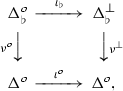(5.12)

where $$\iota$$ is the involution sending $$[n] \in \Delta$$ to the opposite ordinal $$[n]^o$$. Then we also have the functors

\begin{aligned} \tau = \iota _\flat \circ \sigma ^o \circ \iota ^o:\Delta ^o \rightarrow \Delta ^\perp _\flat , \qquad \tau _\dagger = \iota ^o \circ \sigma ^o \circ \iota _\flat ^{-1}:\Delta ^\perp _\flat \rightarrow \Delta ^o, \end{aligned}

and the adjunction map $$\sigma \circ \sigma _\dagger \rightarrow \mathsf{Id}$$ induces a natural map $$\mathsf{Id}\rightarrow \tau \circ \tau _\dagger$$. Explicitly, $$\tau$$ sends [n] to $$\langle [n],n \rangle$$, $$\tau _\dagger$$ sends $$\langle [n],l \rangle$$ to the ordinal $$\{0,\dots ,l\}$$, and the embeddings $$\{0,\dots ,l\} \subset \{0,\dots ,n\} = [n]$$ provide the map $$\mathsf{Id}\rightarrow \tau \circ \tau _\dagger$$. Moreover, for any simplicial set X, we can consider the transpose fibration $$\nu :(\Delta _\flat X)^\perp \rightarrow (\Delta X)^o$$ to the cofibration $$\nu$$, and then $$\tau$$ and $$\tau _\dagger$$ extend to functors

\begin{aligned} \tau :(\Delta X)^o \rightarrow (\Delta _\flat X)^\perp , \qquad \tau _\dagger :(\Delta _\flat X)^\perp \rightarrow (\Delta X)^o, \end{aligned}

and we have a natural map $$\mathsf{Id}\rightarrow \tau \circ \tau _\dagger$$.

### Definition 5.10

A map f in $$(\Delta X)^o$$ is special resp. co-special if $$\iota ^o(f^o)$$ is special resp. co-special in $$\Delta X$$. A map f in $$(\Delta _\flat X)^\perp$$ is special resp. co-special resp. perfect if $$\iota _\flat ^{-1}(f^o)$$ is special resp. co-special resp. perfect in $$\Delta _\flat X$$. A prefibration $$\mathcal {C}$$ over $$(\Delta x)^o$$ is special if $$f^*$$ is an equivalence for any special map f, (4.2) is an isomorphism if both $$f_1$$ and $$f_2$$ are special or co-special, and for any standard square in $$\Delta X$$ with $$f=i_1 \circ p = i \circ p_1$$, the maps (4.2) provide isomorphisms $$\iota (p_1^o)^* \circ \iota (i^o)^* \cong \iota (f^o)^* \cong \iota (i^o_1)^* \circ \iota (p^o)^*$$. It is normalized if in addition, (4.2) is an isomorphism as soon as $$\pi (f_2)$$ is injective in $$\Delta ^o$$. A prefibration $$\mathcal {C}$$ over $$(\Delta _\flat X)^\perp$$ is special if $$f^*$$ is an equivalence for any special f, and (4.2) is an isomorphism as soon as $$f_1$$ is special, or $$f_2$$ is co-special, or $$f_2$$ is special, $$f_1$$ is co-special, and $$f_1 \circ f_2$$ is perfect. As in Definition 5.1, a section of a special prefibration is special if it is cartesian along all special maps, and $${\text {Sec}}_+ \subset {\text {Sec}}$$ denotes the full subcategory spanned by special sections.

Then by the same argument as in Lemma 5.9, the pullback operations $$\tau ^*$$, $$\tau _\dagger ^*$$ send special prefibrations to special prefibrations. Moreover, the map $$a_\dagger :\mathsf{Id}\rightarrow \tau \circ \tau _\dagger$$ is pointwise special, thus compatible with any special prefibration $$\mathcal {C}$$ over $$(\Delta _\flat X)^\perp$$, and strictly compatible if we restrict to the subcategory spanned by special maps. Therefore as in Lemma 5.9, $$a_\dagger ^*:\tau ^*\tau _\dagger ^*\mathcal {C}\rightarrow \mathcal {C}$$ is an equivalence for any special $$\mathcal {C}$$, and this equivalence identifies the categories of special sections.

### Proposition 5.11

Assume given a simplicial set X, and consider its category of simplices $$\Delta X$$. Then for any special prefibration $$\mathcal {C}$$ over $$\Delta X$$, the functor $$\sigma _\dagger ^*\mathcal {C}\rightarrow \Delta X$$ is a cofibration, the functor $$\sigma _\dagger ^*\mathcal {C}\rightarrow \Delta _\flat X$$ is cocartesian over $$\Delta X$$, the transpose functor $$(\sigma _\dagger ^*\mathcal {C})^\perp \rightarrow (\Delta _\flat X)^\perp$$ is a special prefibration, and

\begin{aligned} \mathcal {C}^\flat = \tau ^*\left( \sigma _\dagger ^*\mathcal {C}\right) ^\perp \end{aligned}
(5.13)

is a special prefibration over $$(\Delta X)^o$$. For any special prefibration $$\mathcal {C}'$$ over $$(\Delta X)^o$$, the functor $$\tau _\dagger ^*\mathcal {C}' \rightarrow (\Delta X)^o$$ is a fibration, the functor $$\tau _\dagger ^*\mathcal {C}' \rightarrow (\Delta _\flat X)^\perp$$ is cartesian over $$(\Delta X)^o$$, the transpose functor $$(\tau _\dagger ^*\mathcal {C}')_\perp \rightarrow ((\Delta _\flat X)^\perp )_\perp \cong \Delta _\flat X$$ is a special prefibration, and

\begin{aligned} \mathcal {C}_\flat = \sigma ^*\left( \tau _\dagger ^*\mathcal {C}'\right) _\perp \end{aligned}
(5.14)

is a special prefibration over $$\Delta X$$. Moreover, we have natural equivalences $$(\mathcal {C}^\flat )_\flat \cong \mathcal {C}$$, $$(\mathcal {C}'_\flat )^\flat \cong \mathcal {C}$$, and

\begin{aligned} \begin{aligned} {\text {Sec}}_+(\Delta X,\mathcal {C})&\cong {\text {Sec}}_+((\Delta X)^o,\mathcal {C}^\flat ), \\ {\text {Sec}}_+(\Delta X,\mathcal {C}'_\flat )&\cong {\text {Sec}}_+((\Delta X)^o,\mathcal {C}'), \end{aligned} \end{aligned}
(5.15)

and $$\mathcal {C}$$ is normalized if and only if $$\mathcal {C}^\flat$$ is normalized.

### Proof

For the first claim, note that since $$\mathcal {C}$$ is special, $$\sigma _\dagger ^*\mathcal {C}$$ is special by Lemma 5.9. Then $$\sigma _\dagger ^*\mathcal {C}\rightarrow \Delta X$$ is a precofibration by Definition 5.8 and Lemma 4.12, and by Definition 5.1 (ii), it is actually a cofibration. Thus the transpose functor $$(\sigma ^*_\dagger \mathcal {C})^\perp \rightarrow (\Delta _\flat X)^\perp$$ is well-defined. It is obviously a prefibration, with the transition functor corresponding to a map represented by a diagram (4.20) given by $$v^* \circ c_!$$, and the conditions of Definition 5.10 reduce to the corresponding conditions for $$\sigma _\dagger ^*\mathcal {C}$$. Thus $$(\sigma _\dagger ^*\mathcal {C})^\perp$$ is special, and then so is its restriction $$\mathcal {C}^\flat$$.

Dually, $$\tau _\dagger ^*\mathcal {C}' \rightarrow (\Delta X)^o$$ is a prefibration by virtue of Definition 5.1 (ii) and Lemma 4.11, and Definition 5.1 (ii) then shows that it is actually a fibration, and $$\tau _\dagger ^*\mathcal {C}' \rightarrow (\Delta _\flat X)^\perp$$ is cartesian. Then $$(\tau _\dagger ^*\mathcal {C}')_\perp$$ is well-defined, and again, it is obviously a prefibration with transition functors $$c_! \circ v^*$$. The conditions of Definition 5.8 reduce to those of Definition 5.10, so that the prefibration is special, and so is its restriction $$\mathcal {C}_\flat$$.

Finally, the equivalences $$(\mathcal {C}^\flat )_\flat \cong \mathcal {C}$$, $$(\mathcal {C}'_\flat )^\flat \cong \mathcal {C}$$ immediately follow from (4.21), and the equivalences (5.15) then follows from Lemma 5.9 and its counterpart for $$(\Delta X)^o$$. $$\square$$

### Definition 5.12

For any special prefibration $$\mathcal {C}$$ over $$\Delta X$$ resp. $$(\Delta X)^o$$, the special prefibration $$\mathcal {C}^\flat$$ resp. $$\mathcal {C}_\flat$$ of (5.13) resp. (5.14) is called the reflection of the prefibration $$\mathcal {C}$$. For any special section $$E \in {\text {Sec}}_+(\Delta X,\mathcal {C})$$ resp. $$E \in {\text {Sec}}_+((\Delta X)^o,\mathcal {C})$$, its reflection $$E^\flat$$ resp. $$E_\flat$$ is the corresponding special section of $$\mathcal {C}^\flat$$ resp. $$\mathcal {C}_\flat$$.

One immediate application of reflections is a version of the identification (4.11) relative over $$\Delta X$$. Namely, let $$\Delta ^\flat _\flat \subset \Delta ^\flat \times _\Delta \Delta _\flat$$ be the category of triples $$\langle [n],p,q \rangle$$, $$[n] \in \Delta$$, $$p,q \in [n]$$, $$p \le q$$, with maps from $$\langle [n],p,q \rangle$$ to $$\langle [n'],p',q' \rangle$$ given by maps $$f:[n] \rightarrow [n']$$ such that $$f(p) \ge p'$$ and $$f(q) \le q'$$. The forgetful functor $$\nu :\Delta ^\flat _\flat \rightarrow \Delta$$ is a cofibration, with the fully faithful left-adjoint functor $$\varepsilon :\Delta \rightarrow \Delta ^\flat _\flat$$ sending [n] to $$\langle [n],0,n \rangle$$. Moreover, sending $$\langle [n],p,q \rangle$$ to $$\langle [n],p \rangle$$ resp. $$\langle [n],q \rangle$$ gives a projection from $$\Delta ^\flat _\flat$$ to the category $$\Delta _\flat$$ resp. $$\Delta ^\flat \cong (\Delta ^\perp _\flat )^o$$, and composing these projections with $$\sigma _\dagger$$ resp. $$\tau _\dagger ^o$$, we obtain two projections $$\widetilde{\sigma },\widetilde{\tau }:\Delta ^\flat _\flat \rightarrow \Delta$$.

Now denote $$\Delta ^\flat _\flat X = \Delta ^\flat _\flat \times _\Delta \Delta X$$. By definition, the fibers of the projections $$\nu :\Delta _\flat X \rightarrow \Delta X$$, $$\nu ^\perp :\Delta ^\perp _\flat \rightarrow \Delta X$$ over an object $$\langle [n],x \rangle \in \Delta X$$ are both canonically identified with $$[n]^o$$, and the prefibrations $$\sigma _\dagger ^*\mathcal {C}$$, $$\tau _\dagger ^*\mathcal {C}^\flat$$ restrict to the same prefibration on this fiber $$[n]^o$$. The fiber of the cofibration $$\nu :\Delta ^\flat _\flat X \rightarrow \Delta X$$ over $$\langle [n],x \rangle$$ is then canonically identified with the twisted arrow category $$\mathsf{A}([n]^o)$$, and for any sections $$A,B \in {\text {Sec}}_+(\Delta X,\mathcal {C})$$, we can form the functor

\begin{aligned} {\mathcal {H}{} { om}}\left( \tau _\dagger ^*A^\flat ,\sigma _\dagger ^*B\right) :\mathsf{A}([n]^o) \cong \left( \Delta ^\flat _\flat X\right) _{\langle [n],x \rangle } \rightarrow {\text {Sets}}. \end{aligned}
(5.16)

Moreover, for any $$f:\langle [n],p,q,x \rangle \rightarrow \langle [n'],p',q',x' \rangle$$ in $$\Delta ^\flat _\flat X$$, we have a map

\begin{aligned} \begin{aligned} {\text {Hom}}\left( \tau _\dagger ^*A^\flat ,\sigma _\dagger ^*B\right) (\langle [n],p,q,x \rangle )&\rightarrow {\text {Hom}}\left( \tau _\dagger ^*A^\flat ,\sigma _\dagger ^*B\right) (\langle [n'],p',q',x' \rangle ),\\ g&\mapsto B(\widetilde{\sigma }(f)) \circ g \circ A^\flat (\widetilde{\tau }^o(f)), \end{aligned} \end{aligned}
(5.17)

so that the functors (5.16) for different objects $$\langle [n],x \rangle$$ patch together to a single functor $${\mathcal {H}{} { om}}(\tau _\dagger ^*A^\flat ,\sigma _\dagger ^*B):\Delta ^\flat _\flat X \rightarrow {\text {Sets}}$$. By restriction, we obtain a natural functor $${\mathcal {H}{} { om}}(A^\flat ,B) = \varepsilon ^*{\mathcal {H}{} { om}}(\tau _\dagger ^*A^\flat ,\sigma _\dagger ^*B)$$ from $$\Delta X$$ to sets.

### Lemma 5.13

For any $$A,B \in {\text {Sec}}_+(\Delta X,\mathcal {C})$$, we have

\begin{aligned} {\text {Hom}}(A,B) \cong \mathsf{lim}_{\Delta ^\flat _\flat X}{\mathcal {H}{} { om}}(\tau _\dagger ^*A^\flat ,\sigma _\dagger ^*B) \cong \mathsf{lim}_{\Delta X}{\mathcal {H}{} { om}}(A^\flat ,B). \end{aligned}
(5.18)

### Proof

Since $$\varepsilon :\Delta X \rightarrow \Delta ^\flat _\flat X$$ admits a right-adjoint functor, $$\mathsf{lim}_{\Delta X}\varepsilon ^*F \cong \mathsf{lim}_{\Delta ^\flat _\flat X}F$$ for any $$F:\Delta ^\flat _\flat X \rightarrow {\text {Sets}}$$, so we have the second identification in (5.18). For the first one, note that by (4.11), an element in the limit defines a map $$\tau _\dagger ^*A^\flat |_{\langle [n],x \rangle } = \sigma _\dagger ^*A|_{\langle [n],x \rangle } \rightarrow \sigma _\dagger ^*B|_{\langle [n],x \rangle }$$, and these maps patch together into a single map $$\sigma _\dagger ^*A \rightarrow \sigma _\dagger ^*B$$ if and only if they are compatible with the maps (5.17). $$\square$$

## Simplicial replacements II

We can now use the technology of special prefibrations to describe the gluing constructions of Sect. 4.5 purely in terms of sections. First, we do it in the case of the trivial base category $$I=\mathsf{pt}$$ and non-normalized lax functors. In Sect. 6.1, we associate a special prefibration over $$\Delta$$ to a comonad and construct a functor from the category of coalgebras over a comonad to the category of sections of the prefibration (this is Lemma 6.4). Then in Sect. 6.2, we show that this is in fact a one-to-one correspondence between comonads and special prefibrations over $$\Delta$$ (Proposition 6.7) that identifies special sections and coalgebra over the comonad (Lemma 6.6). In Sect. 6.3, we turn to normalized lax functors—that is, precofibrations—over an arbitrary I. The main result is Proposition 6.11 that establishes a one-to-one correspondence between precofibrations over I and normalized special fibrations over its simplicial replacement $$\Delta I$$, and shows that this correspondence also identifies cosections of the precofibration and special sections of the special prefibration. Finally, in Section 6.6, we illustrate our abstract canonical construction by explicit expressions in terms of the lax functors.

### Barycentric expansions

We start with comonads. We will show that coalgebras over a comonad naturally appear as sections of a special prefibration over the category $$\Delta$$.

First, assume given a functor $$F:C_0 \rightarrow \mathcal {C}_1$$ between two categories, and for any $$[n] \in \Delta$$, define a category $$F^{[n]}$$ by the cartesian square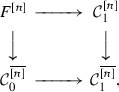(6.1)

where we treat the totally ordered set $$[n] = \{0,\dots ,n\}$$ as a small category in the usual way, and we denote by $$\overline{[n]} \subset [n]$$ the same set considered as a discrete category. Explicitly, an object in $$F^{[n]}$$ is given by a pair $$\langle \kappa ,\{c_\cdot \}\rangle$$ of a functor $$\kappa :[n] \rightarrow \mathcal {C}_1$$ an a collection of objects $$c_l \in \mathcal {C}_0$$, $$0 \le l \le n$$, equipped with isomorphisms $$\alpha _l:F(c_l) \cong \kappa (l)$$. In particular, we have $$F^{} \cong \mathcal {C}_0$$.

For any map $$f:[n_1] \rightarrow [n_2]$$ in $$\Delta$$, we have the obvious pullback functor $$f^*:F^{[n_2]} \rightarrow F^{[n_1]}$$, and these are compatible with compositions, so that the categories $$F^{[n]}$$ form a fibration $$S(F) \rightarrow \Delta$$ with fibers $$S(F)_{[n]} = F^{[n]}$$ and transition functors $$f^*$$.

Now recall that we have the subcategory $$\Delta _+ \subset \Delta$$, and by definition, $$ \in \Delta _+$$ is the initial object, so that for any $$[n] \in \Delta _+ \subset \Delta$$ we have a unique map $$s: \rightarrow [n]$$.

### Lemma 6.1

Assume that the functor F has a left-adjoint $$F_\dagger :\mathcal {C}_1 \rightarrow \mathcal {C}_0$$. Then for any $$[n] \in \Delta _+ \subset \Delta$$, the pullback functor $$s^*:F^{[n]} \rightarrow F^{}$$ admits a fully faithful left-adjoint $$s_!$$. Moreover, an object $$\langle \kappa ,\{c_\cdot \}\rangle \in F^{[n]}$$ lies in the essential image of the functor $$s_!$$ iff for any i, $$0 \le i < n$$, the map $$F_\dagger (\kappa (i)) \rightarrow c_{i+1}$$ adjoint to the map $$\kappa (i) \rightarrow \kappa (i+1) \cong F(c_{i+1})$$ is an isomorphism.

### Proof

For any $$[n] \in \Delta$$, denote by $$s_n:[n] \rightarrow [n+1]$$ the embedding onto the initial segment of the ordinal $$[n+1]$$ (that is, $$s_n(l) = l$$, $$0 \le l \le n$$). Then it is immediately obvious that $$s_n^*$$ does have a left-adjoint $$s_{n!}$$: it extends a pair $$\langle \kappa ,\{c_\cdot \}\rangle$$ by setting $$c_{n+1} = F_\dagger (\kappa (n))$$, $$\kappa (n+1)=F(c_{n+1})$$, and letting the map $$\kappa (n) \rightarrow \kappa (n+1)=F(c_{n+1})$$ be adjoint to the isomorphism $$F_\dagger (\kappa (n)) \cong c_{n+1}$$. Since $$s_n^* \circ s_{n!} \cong \mathsf{Id}$$ by construction, $$s_{n!}$$ is fully faithful, and since the map $$s: \rightarrow [n]$$ is the composition of embeddings $$s_l$$, $$0 \le l < n$$, it has a full faithful left-adjoint by induction, and the same induction describes its essential image. $$\square$$

Now assume given a comonad $$\Phi$$ on a category $$\mathcal {C}$$, and let $$F:\mathcal {C}\rightarrow {\text {Coalg}}(\Phi ,\mathcal {C})$$ be the free coalgebra functor. By definition, it does have a left-adjoint given by the forgetful functor, so we are within the framework of Lemma 5.4.

### Definition 6.2

The barycentric expansion $$S(\mathcal {C},\Phi )$$ of the comonad $$\Phi$$ is the full subcategory $$S(\mathcal {C},\Phi ) \subset S(F)$$ spanned by the essential images of the fully faithful embeddings $$s_!:\mathcal {C}\cong F^{} \rightarrow F^{[n]}$$ of Lemma 6.1.

### Remark 6.3

Note that for any functor $$\widetilde{F}:\mathcal {C}\rightarrow \widetilde{\mathcal {C}}$$ that has a left-adjoint $$\widetilde{F}_\dagger$$ such that $$\Phi \cong \widetilde{F}_\dagger \circ \widetilde{F}$$ as a comonad, and for any $$[n] \in \Delta$$, the comparison functor (4.33) induces a functor $$\widetilde{F}^{[n]} \rightarrow F^{[n]}$$. Moreover, it is immediately obvious from the definitions that this functor is an equivalence that identifies the essential images of the fully faithful embeddings $$s_!$$ of Lemma 5.4. Therefore to define the barycentric subdivision, we can replace $${\text {Coalg}}(\Phi ,\mathcal {C})$$ with $$\widetilde{\mathcal {C}}$$ and F with $$\widetilde{F}$$ without changing the result.

By Lemma 6.1, the subcategory $$S(\mathcal {C},\Phi ) \subset S(F)$$ is as in Example 4.9, so that the projection $$S(\mathcal {C},\Phi ) \rightarrow \Delta$$ is a prefibration. If $$\Phi = \mathsf{Id}$$ is the trivial comonad, then $$S(\mathcal {C},\Phi ) \cong \mathcal {C}\times \Delta$$ is the trivial prefibration with fibers $$\mathcal {C}= S(\mathcal {C},\Phi )_{}$$. In the general case, the fibers are the same, but the transition functors are non-trivial and depend on $$\Phi$$.

Moreover, assume given two categories $$\mathcal {C}$$, $$\mathcal {C}'$$ equipped with comonads $$\Phi$$, $$\Phi '$$ generated by functors $$F:\mathcal {C}\rightarrow \widetilde{\mathcal {C}}$$, $$F':\mathcal {C}' \rightarrow \widetilde{\mathcal {C}}'$$, as in Remark 6.3. Assume also given functors $$\gamma :\mathcal {C}\rightarrow \mathcal {C}'$$, $$\widetilde{\gamma }:\widetilde{\mathcal {C}} \rightarrow \widetilde{\mathcal {C}'}$$ equipped with a pair of adjoint isomorphisms

\begin{aligned} F' \circ \gamma \cong \widetilde{\gamma } \circ F, \qquad F'_\dagger \circ \widetilde{\gamma } \cong \gamma \circ F_\dagger . \end{aligned}

Then for any $$[n] \in \Delta$$, $$\gamma$$ and $$\widetilde{\gamma }$$ induce a functor $$S(F)_{[n]} \rightarrow S(F')_{[n]}$$, these functors are compatible with pullbacks, and send $$S(\mathcal {C},\Phi )_{[n]} \subset S(F)_{[n]}$$ into $$S(\mathcal {C}',\Phi ') \subset S(F')_{[n]}$$. Therefore we obtain a natural functor

\begin{aligned} S(\gamma ):S(\mathcal {C},\Phi ) \rightarrow S(\mathcal {C}',\Phi ') \end{aligned}
(6.2)

cartesian over $$\Delta$$.

As an application of this functoriality, let $$F:\mathcal {C}\rightarrow \widetilde{\mathcal {C}}$$, $$F_\dagger :\widetilde{\mathcal {C}} \rightarrow \mathcal {C}$$ be a pair of adjoint functors generating a comonad $$\Phi$$ on a category $$\mathcal {C}$$, and consider the right comma-category $$\mathsf{R}(F)$$ of the functor F with the projections (3.2). By adjunction, we have $$\mathsf{R}(F) \cong \mathsf{L}(F_\dagger )$$, and we have a natural functor

\begin{aligned} F':\mathsf{L}(F_\dagger ) \rightarrow \widetilde{\mathcal {C}}^{} \end{aligned}

sending a triple $$\langle c',c,\alpha \rangle$$ to the map $$\alpha _\dagger :c' \rightarrow F(c)$$ adjoint to $$\alpha :F_\dagger (c') \rightarrow c$$. This functor has a left-adjoint $$F'_\dagger$$, thus generates a comonad $$\Phi '$$ on $$\mathsf{R}(F)$$, and we have pairs of adjoint isomorphisms

\begin{aligned} \widetilde{\tau } \circ F' \cong F \circ \tau , \quad \tau \circ F'_\dagger \cong F_\dagger \circ \widetilde{\tau }, \qquad \widetilde{\sigma } \circ F' \cong \sigma , \quad \sigma \circ F'_\dagger \cong \widetilde{\sigma } \end{aligned}

where $$\widetilde{\sigma } = \mathsf{ev}_0$$ and $$\widetilde{\tau }=\mathsf{ev}_1$$ are evaluations at $$0,1 \in $$. Therefore we have the functors $$S(\tau )$$, $$S(\sigma )$$ of (6.2) cartesian over $$\Delta$$. Moreover, for any $$[n] \in \Delta$$, we have $$S(\sigma )([n]) \cong \sigma$$, and since $$\sigma$$ has a left-adjoint, $$S(\sigma )$$ also has a left-adjoint functor

\begin{aligned} S(\sigma )_\dagger :\widetilde{\mathcal {C}} \times \Delta \cong S(\widetilde{\mathcal {C}},\mathsf{Id}) \rightarrow S(\mathsf{R}(F),\Phi ') \end{aligned}

given by (4.23). We then have the composition functor

\begin{aligned} S(\tau ) \circ S(\sigma )_\dagger :\widetilde{\mathcal {C}} \times \Delta \rightarrow S(\mathcal {C},\Phi ). \end{aligned}
(6.3)

Since every object $$c \in \widetilde{\mathcal {C}}$$ tautologically induces a section of the trivial fibration $$\widetilde{\mathcal {C}} \times \Delta \rightarrow \Delta$$, this induces a functor

\begin{aligned} \widetilde{\mathcal {C}} \rightarrow {\text {Sec}}(\Delta ,S(\mathcal {C},\Phi )). \end{aligned}
(6.4)

In particular, we can take $$\widetilde{\mathcal {C}} = {\text {Coalg}}(\mathcal {C},\Phi )$$. Thus every coalgebra over the comonad $$\Phi$$ generates a section of the barycentric expansion $$S(\mathcal {C},\Phi ) \rightarrow \Delta$$.

### Lemma 6.4

For any comonad $$\Phi$$ on a category $$\mathcal {C}$$, the barycentric expansion $$S(\mathcal {C},\Phi ) \rightarrow \Delta$$ of Definition 6.2 is a special prefibration in the sense of Definition 5.1, and the functor (6.4) induces a functor

\begin{aligned} {\text {Coalg}}(\mathcal {C},\Phi ) \rightarrow {\text {Sec}}_+(\Delta ,S(\mathcal {C},\Phi )) \end{aligned}
(6.5)

from the category of coalgebras over the comonad $$\Phi$$ to the category of special sections of its barycentric expansion $$S(\mathcal {C},\Phi ) \rightarrow \Delta$$.

### Proof

For the first claim, it suffices to check that $$S(\mathcal {C},\Phi )$$ satisfies the conditions of Lemma 5.4, and this immediately follows from Lemma 6.1. For the second claim, it suffices to check that the functor (6.3) is cartesian along special maps, and since $$S(\tau )$$ is cartesian, this amounts to checking that $$S(\sigma )_\dagger$$ is cartesian along special maps. Indeed, by definition the maps (4.5) for this functor are adjoint to the corresponding maps for the cartesian functor $$S(\sigma )$$, and since $$f^*$$ is an equivalence for a special map f, these maps are isomorphisms. $$\square$$

Consider now the adjoint pair of functors $$\rho :\Delta _+ \rightarrow \Delta$$, $$\lambda :\Delta \rightarrow \Delta _+$$ of Example 4.22. As before, denote $$\varphi = \lambda \circ \rho$$, $$\varphi _\dagger =\rho \circ \lambda$$, and let $$a:\mathsf{id}\rightarrow \varphi _\dagger$$, $$a_\dagger :\varphi \rightarrow \mathsf{id}$$ be the adjunction maps. For any simplicial set X with the discrete fibration $$\Delta X \rightarrow \Delta$$, denote $$\Delta _+X = \rho ^*\Delta X$$ and $$\Delta _<X = \varphi _\dagger ^*\Delta X$$. The adjunction map a then induces a functor

\begin{aligned} a_X:\Delta _< X \rightarrow \Delta X, \qquad \langle [n],x \rangle \mapsto \langle [n],a([n])^*x \rangle , \end{aligned}
(6.6)

and the adjunction map $$a_\dagger$$ induces a functor

\begin{aligned} \rho _X:\Delta _+ X \rightarrow \Delta _< X, \qquad \rho _X(\langle [n],x \rangle ) = \langle \rho ([n]),a_\dagger (\rho ([n]))^*x \rangle . \end{aligned}
(6.7)

The tautological embedding $$\lambda :\Delta _+ X \rightarrow \Delta _< X = \lambda ^*\Delta _+ X$$ is left-adjoint to $$\rho _X$$, and the composition $$a_X \circ \rho _X:\Delta _+ X = \rho ^* \Delta X \rightarrow \Delta X$$ is the tautological embedding $$\rho$$. In fact, sending $$\langle [n],x \rangle$$ to $$s^*x$$ gives a projection from $$\Delta _+X$$ to the set X() considered as a discrete category, so that we have natural decompositions

\begin{aligned} \Delta _+ X = \coprod _{x \in X()} (\Delta _+ X)_x, \qquad \Delta _< X = \coprod _{x \in X()} (\Delta _< X)_x, \end{aligned}
(6.8)

and for any $$x \in X()$$, the adjoint functors $$\rho _X$$, $$\lambda$$ induce a pair of adjoint functors

\begin{aligned} \rho _x:(\Delta _+ X)_x \rightarrow (\Delta _<X)_x, \qquad \lambda _x:(\Delta _<X)_x \rightarrow (\Delta _+X)_x. \end{aligned}
(6.9)

### Lemma 6.5

Assume given a special prefibration $$\mathcal {C}$$ over $$\Delta X$$ in the sense of Definition 5.1, and let $$\mathcal {C}' = a_X^*\mathcal {C}$$ be its preimage under the functor (6.6). Then the functor $$\rho _X$$ of (6.7) and the prefibration $$\mathcal {C}'$$ satisfy the assumptions of Lemma 4.18, so that the pullback functor $$\rho _X^*$$ admits a left-adjoint functor $$\lambda ^{\mathcal {C}'}:{\text {Sec}}(\Delta _+X,\rho _X^*\mathcal {C}') \rightarrow {\text {Sec}}(\Delta _<X,\mathcal {C}')$$. Moreover, $$\lambda ^{\mathcal {C}'}$$ sends cartesian sections to special sections, and if $$\mathcal {C}$$ is normalized, then $$\lambda ^{\mathcal {C}'}$$ is fully faithful.

### Proof

The left-adjoint to $$\rho _X$$ is the tautological embedding $$\lambda$$, and the fact that the adjunction map $$a:\mathsf{id}\rightarrow \rho _X \circ \lambda$$ is compatible with $$\mathcal {C}'$$ immediately follows from Definition 5.1 (iii). It also follows from Definition 5.1 that a becomes strictly compatible with $$\mathcal {C}'$$ after restricting to $$\Delta _+X$$, so that $$\lambda ^{\mathcal {C}'}$$ sends cartesian sections to special sections. Moreover, the adjunction map $$\mathsf{Id}\rightarrow \rho _X^* \circ \lambda ^{\mathcal {C}'}$$ is induced by the map $$\alpha (a_\dagger ,a)$$ of (4.2) for $$\mathsf{Id}= a_\dagger \circ a$$, and since $$a_\dagger$$ is surjective, this is an isomorphism if $$\mathcal {C}$$ is normalized. $$\square$$

In particular, we can apply this result to comonads and their barycentric expansions of Sect. 6.1. This corresponds to the case of the trivial simplicial set $$X = \mathsf{pt}$$, so that $$\Delta X = \Delta _< X = \Delta$$, $$\Delta _+ X = \Delta _+$$, $$a_X = \mathsf{id}$$ and $$\rho _X=\rho$$. Assume given a special prefibration $$\pi :\mathcal {C}\rightarrow \Delta$$, and consider its restriction $$\mathcal {C}_+ = \rho ^*\mathcal {C}$$ with respect to the embedding $$\rho :\Delta _+ \rightarrow \Delta$$. By Definition 5.1 (i), (ii), this is a locally constant fibration. Moreover, since $$ \in \Delta _+$$ is the initial object, the embedding $$\mathcal {C}_{} \subset \mathcal {C}_+$$ of the fiber at  has a right-adjoint functor

\begin{aligned} \chi :\mathcal {C}_+ \rightarrow \mathcal {C}_{}, \qquad c \mapsto s^*c, \end{aligned}
(6.10)

where $$s: \rightarrow [n]$$ is the unique map, and the product $$\chi \times \pi :\mathcal {C}_+ \rightarrow \mathcal {C}_{} \times \Delta _+$$ is an equivalence of categories. Thus $$\mathcal {C}_+ \rightarrow \Delta _+$$ is a trivial fibration, and evaluation at $$ \in \Delta _+$$ induces an equivalence

\begin{aligned} {\text {Sec}}_\natural (\Delta _+,\mathcal {C}_+) \cong \mathcal {C}_{}. \end{aligned}
(6.11)

Lemma 6.5 provides a comonad $$\Phi = \lambda ^{\mathcal {C}} \circ \rho$$ on the category $${\text {Sec}}(\Delta _+,\mathcal {C}_+)$$ that restricts to a comonad

\begin{aligned} \Phi :{\text {Sec}}_\natural (\Delta _+,\mathcal {C}_+) \rightarrow {\text {Sec}}_\natural (\Delta _+,\mathcal {C}_+) \end{aligned}
(6.12)

on $${\text {Sec}}_\natural (\Delta _+,\mathcal {C}_+) \cong \mathcal {C}_{}$$. Since $$\Delta$$ is the Kleisli category of the comonad $$\varphi$$ on $$\Delta _+$$, Lemma 4.24 then shows that we have a natural equivalence

\begin{aligned} {\text {Sec}}_+(\Delta ,\mathcal {C}) \cong {\text {Coalg}}(\mathcal {C}_{},\Phi ). \end{aligned}
(6.13)

In particular, if $$\mathcal {C}$$ is normalized in the sense of Definition 5.1 (iv), then $$\Phi = \mathsf{Id}$$ by Lemma 6.5, and $$\mathcal {C}$$ is canonically trivial (that is, we have $$\mathcal {C}\cong \mathcal {C}_{} \times \Delta$$).

### Lemma 6.6

For any comonad $$\Phi '$$ on a category $$\mathcal {C}$$, with barycentric expansion $$S(\mathcal {C},\Phi ')$$, the comonad $$\Phi$$ of (6.12) on $$\mathcal {C}= S(\mathcal {C},\Phi ')_{}$$ is canonically isomorphic to $$\Phi '$$, and the functor (6.5) is an equivalence of categories inverse to (6.13).

### Proof

Denote by $$F':\mathcal {C}\rightarrow {\text {Coalg}}(\mathcal {C},\Phi ')$$, $$F:\mathcal {C}\rightarrow {\text {Coalg}}(\mathcal {C},\Phi )$$ the free coalgebra functors, with adjoint forgetful functors $$F'_\dagger$$, $$F_\dagger$$, and consider the composition

\begin{aligned} K:{\text {Coalg}}(\mathcal {C},\Phi ') \rightarrow {\text {Coalg}}(\mathcal {C},\Phi ) \end{aligned}

of the functors (6.5) and (6.13). In terms of the equivalence (6.13), the forgetful functor $$F_\dagger$$ is given by evaluation at $$ \in \Delta$$, and since the functor (6.3) by definition restricts to $$F'_\dagger$$ at , we have $$F'_\dagger \cong F_\dagger \circ K$$. By adjunction, we then have a map $$K \circ F' \rightarrow F$$, and this map becomes an isomorphism after applying $$F_\dagger$$. Since $$F_\dagger$$ is conservative, it was an isomorphism to begin with. This gives an isomorphism of comonads $$\Phi ' \cong \Phi$$, and K is the associated equivalence of categories. Since (6.13) is an equivalence, (6.5) is an equivalence as well. $$\square$$

### Proposition 6.7

Assume given a special prefibration $$\pi :\mathcal {C}\rightarrow \Delta$$, and let $$\Phi$$ be the comonad (6.12). Then we have a natural equivalence

\begin{aligned} \mathcal {C}\cong S(\mathcal {C}_{},\Phi ) \end{aligned}
(6.14)

of prefibrations over $$\Delta$$.

### Proof

To simplify notation, denote $$\mathcal {C}' = {\text {Sec}}_+(\Delta ,\mathcal {C})$$. Since $$\mathcal {C}_+ = \rho ^*\mathcal {C}\rightarrow \Delta _+$$ is a trivial fibration, evaluation $$\mathsf{ev}_{[n]}$$ at any $$[n] \in \Delta$$ restricts to an equivalence $${\text {Sec}}_\natural (\Delta _+,\mathcal {C}_+) \cong \mathcal {C}_{[n]}$$, as in (6.11), and since the restriction functor $$\mathcal {C}' \rightarrow {\text {Sec}}_\natural (\Delta _+,\mathcal {C}_+)$$ admits a right-adjoint functor of Lemma 6.5, $$\mathsf{ev}_{[n]}$$ also admits a right-adjoint functor $$\chi _\natural :\mathcal {C}_{[n]} \rightarrow \mathcal {C}'$$. In terms of the equivalence (6.13), we have

\begin{aligned} \chi _\natural (c) \cong F(\chi (c)), \qquad c \in \mathcal {C}_{[n]}, \end{aligned}
(6.15)

where $$\chi$$ is the functor (6.10), and $$F:\mathcal {C}_{} \rightarrow {\text {Coalg}}(\mathcal {C}_{},\Phi ) \cong \mathcal {C}'$$ is the free coalgebra functor.

Consider now the canonical embedding $$\mathcal {C}\subset B(\mathcal {C})$$ of Lemma 5.7. Then evaluation functors $$\mathsf{ev}_{[n]}$$ together define a functor

\begin{aligned} \mathsf{ev}:\mathcal {C}' \times \Delta _\flat \rightarrow \sigma _\dagger ^*\mathcal {C}\end{aligned}
(6.16)

over $$\Delta _\flat$$, and since for any special map $$f:[n] \rightarrow [n']$$, we have $$\mathsf{ev}_{[n]} \cong f^* \circ \mathsf{ev}_{[n']}$$ and $$f_! \circ \mathsf{ev}_{[n]} \cong \mathsf{ev}_{[n']}$$, the functor (6.16) is cocartesian over $$\Delta$$. Then (4.30) provides a functor

\begin{aligned} \mathsf{ev}^\perp :B(\mathcal {C}) = \nu ^\perp \sigma _\dagger \mathcal {C}\rightarrow \nu ^\perp (\mathcal {C}' \times \Delta _\flat ). \end{aligned}

The target of this functor is by definition the simplicial expansion $$\Delta _\tau \mathcal {C}'$$, $$\tau$$ the class of all maps, and its fiber over $$[n] \in \Delta$$ is the category of functors from [n] to $$\mathcal {C}'$$. The source is given by (5.11), and for any $$l \in [n]$$ and $$\sigma \in B(\mathcal {C})_{[n]}$$, we have $$\mathsf{ev}^\perp (\sigma )(l) \cong \chi _\natural (\sigma (l))$$. Then $$\mathsf{ev}^\dagger (\sigma )$$ with the collection $$\{\chi (\sigma (l))\}$$ and isomorphisms (6.15) is a well-defined object in the category $$F^{[n]}$$ for the free coalgebra functor $$F:\mathcal {C}_{} \rightarrow \mathcal {C}'$$, so that we obtain a functor $$B(\mathcal {C})_{[n]} \rightarrow F^{[n]}$$. Comparing (5.11) and the definition of the category $$F^{[n]}$$, we see that this functor is in fact an equivalence. Moreover, these equivalences for different [n] are compatible with maps $$f:[n] \rightarrow [n']$$, and taken together, they define an equivalence $$B(\mathcal {C}) \cong S(F)$$ of fibrations over $$\Delta$$. By Lemma 5.7 and Definition 6.2, this induces an equivalence (6.14). $$\square$$

### Cosections

Now assume given a small category I, with its simplicial replacement $$\Delta I = \Delta NI$$, and a precofibration $$\mathcal {C}\rightarrow I$$. Let $$\widetilde{S}(\mathcal {C}) = \Delta _v\mathcal {C}$$, and let $$S(\mathcal {C}) = \Delta ^c_v \mathcal {C}\subset \widetilde{S}(\mathcal {C})$$, where c resp. v are the classes of cocartesian resp. vertical maps in $$\mathcal {C}$$. The precofibration $$\mathcal {C}\rightarrow I$$ then induces a functor $$\widetilde{S}(\mathcal {C}) \rightarrow \Delta I$$ that restricts to a functor $$S(\mathcal {C}) \rightarrow \Delta I$$.

### Lemma 6.8

The functor $$S(\mathcal {C}) \rightarrow \Delta I$$ is a normalized special prefibration in the sense of Definition 5.1.

### Proof

Since the class of all maps is closed under compositions, the projection $$\widetilde{\mathcal {C}} \rightarrow \Delta$$ is a fibration, and since $$\Delta I \rightarrow I$$ is a discrete fibration, $$\widetilde{S}(\mathcal {C}) \rightarrow \Delta I$$ is a fibration as well (a cartesian lifting of a map is given by the cartesian lifting of its image in $$\Delta$$). By definition, we have a full embedding $$S(\mathcal {C}) \subset \widetilde{S}(\mathcal {C})$$, and it immediately follows from the definition of a cocartesian map that the fibration $$\widetilde{S}(\mathcal {C}) \rightarrow \Delta I$$ generating of Definition 5.3, and $$S(\mathcal {C}) \subset \widetilde{S}(\mathcal {C})$$ is the associated special prefibration. Finally, since identity maps are always cocartesian, for any surjective map $$f:[n'] \rightarrow [n]$$ and $$\kappa :[n] \rightarrow I$$, the corresponding functor $$f^*$$ of the fibration $$\widetilde{S}(\mathcal {C})$$ sends $$S(\mathcal {C})_{[n,\kappa ]} \subset \widetilde{S}(\mathcal {C})_{[n,\kappa ]}$$ into $$S(\mathcal {C})_{[n',f^*\kappa ]} \subset \widetilde{S}(\mathcal {C})_{[n',f^*\kappa ]}$$. Therefore the special prefibration $$S(\mathcal {C}) \rightarrow \Delta I$$ is normalized. $$\square$$

### Definition 6.9

The special prefibration $$S(\mathcal {C}) \rightarrow \Delta I$$ is called the barycentric expansion of the precofibration $$\mathcal {C}\rightarrow I$$.

Conversely, to recover a precofibration over I from a special prefibration over $$\Delta I$$, consider the functor $$\xi$$ of (5.4) and its left comma-category $$\mathsf{L}(\xi )$$, with projections $$\sigma$$, $$\tau$$ of (3.2). Then $$\tau$$ is a cofibration by Example 4.4. Moreover, denote $$\Delta _+I = \Delta _+NI$$, $$\Delta _<I = \Delta _< NI$$, and let $$(\Delta _+ I)_i$$, $$(\Delta _< I)_i$$, $$i \in NI()$$ be the terms of the decompositions (6.8). Then NI() is by definition the set of its objects of I, and for any object $$i \in I$$, we have an obvious identification

\begin{aligned} \mathsf{L}(\xi )_i \cong (\Delta _< NI)_i, \end{aligned}
(6.17)

where $$\mathsf{L}(\xi )_i$$ is the fiber of the cofibration $$\tau$$. The projection $$\sigma$$ then restricts to a functor

\begin{aligned} \sigma _i:\mathsf{L}(\xi )_i \rightarrow \Delta I, \end{aligned}
(6.18)

and in terms of the identification (6.17), this functor is induced by the projection (6.6). Moreover, any $$f:i' \rightarrow i$$ in I gives a morphism $$f^o$$ in $$I^o$$, and the corresponding transition functor $$f^o_!$$ of the cofibration $$\tau$$ fits into a commutative diagram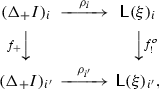(6.19)

where the horizontal lines are the functors (6.9), and $$f_+$$ takes a pair $$\langle [n],\kappa \rangle \in (\Delta _+)_i$$, adds a new initial element o to [n], and extends $$\kappa$$ by setting $$\kappa (o)=i'$$.

Now assume given a normalized special prefibration $$\mathcal {C}$$ over $$\Delta I$$. Then for any $$i \in I$$, its preimage $$\sigma _i^*\mathcal {C}$$ with respect to the functor (6.18) is a normalized special prefibration on $$\mathsf{L}(\xi )_i$$, and Lemma 6.5 provides a fully faithful embedding

\begin{aligned} \lambda ^\mathcal {C}_i = \lambda ^{\sigma _i^*\mathcal {C}}:{\text {Sec}}_\natural ((\Delta _+NI)_i,\rho _i^*\sigma _i^*\mathcal {C}) \rightarrow {\text {Sec}}_+(\mathsf{L}(\xi )_i,\sigma _i^*\mathcal {C}). \end{aligned}
(6.20)

Moreover, since $$\sigma$$ inverts maps cocartesian with respect to $$\tau$$, the transition functors of the cofibration $$\tau$$ commute with the projections $$\sigma _i$$. We can therefore define the category $$\widetilde{R}(\mathcal {C})$$ as follows:

1. (i)

objects are pairs $$\langle i,s \rangle$$ of an object $$i \in I$$ and a special spection $$s \in {\text {Sec}}_+(\mathsf{L}(\xi )_i,\sigma _i^*\mathcal {C})$$,

2. (ii)

morphisms from $$\langle i,s \rangle$$ to $$\langle i',s' \rangle$$ are pairs $$\langle f,\alpha \rangle$$ of a morphism $$f:i \rightarrow i'$$ and a morphism $$(f^o_!)^*s \rightarrow s'$$.

With this definition, the natural forgetful functor $$\widetilde{R}(\mathcal {C}) \rightarrow I$$ is a cofibration, with fibers

\begin{aligned} \widetilde{R}(\mathcal {C})_i \cong {\text {Sec}}_+(\mathsf{L}(\xi )_i,\sigma _i^*\mathcal {C}) \end{aligned}

and transition functors $$(f^o_!)^*$$.

### Definition 6.10

The reduction $$R(\mathcal {C})$$ of the normalized special prefibration $$\mathcal {C}$$ is the full subcategory $$R(\mathcal {C}) \subset \widetilde{R}(\mathcal {C})$$ spanned by the essential images of the fully faithful functors (6.20).

Note that by Lemma 6.5, the functors $$\lambda ^\mathcal {C}_i$$ of (6.20) are left-adjoint to the pullback functors $$\rho _i^*$$, so that as in Example 4.9, the projection $$R(\mathcal {C}) \rightarrow I$$ induced by the cofibration $$\widetilde{R}(\mathcal {C}) \rightarrow I$$ is a precofibration.

### Proposition 6.11

Assume given a small category I. Then for any precofibration $$\mathcal {C}\rightarrow I$$, the reduction $$R(S(\mathcal {C}))$$ of its barycentric expansion $$S(\mathcal {C})$$ of Definition 6.9 is naturally equivalent to $$\mathcal {C}$$, and for any normalized special prefibration $$\mathcal {C}' \rightarrow \Delta I$$, the barycentric expansion $$S(R(\mathcal {C}'))$$ of its reduction $$R(\mathcal {C}')$$ is naturally equivalent to $$\mathcal {C}'$$. Moreover, we have natural equivalences of categories

\begin{aligned} {\text {Rec}}(I,\mathcal {C}) \cong {\text {Sec}}_+(\Delta I,S(\mathcal {C})), \qquad {\text {Rec}}(I,R(\mathcal {C}')) \cong {\text {Sec}}_+(\Delta I,\mathcal {C}'), \end{aligned}
(6.21)

where $${\text {Sec}}_+$$ are the categories of special sections of Definition 5.1.

### Proof

For the first claim, consider the cofibration $$\nu :\Delta ^\flat \rightarrow \Delta$$ of Example 4.7, say that a map $$f:\langle [n],l \rangle \rightarrow \langle [n'],l' \rangle$$ is vertical if it is vertical over $$\Delta$$, and say that f is horizontal if $$l=l'$$ and $$f(j)=j$$, $$0 \le j \le l$$. Let $$\Delta _+^\flat = \Delta _+ \times _\Delta \Delta ^\flat$$, and note that the embedding $$\lambda :\Delta \rightarrow \Delta _+$$ extends to an embedding

\begin{aligned} \lambda ^\flat :\Delta ^\flat \rightarrow \Delta _+^\flat \end{aligned}
(6.22)

that adds a new initial element to an ordinal [n], but keeps the same distinguished element l. Let $$\Delta ^\flat I = \Delta I \times _\Delta \Delta ^\flat$$, and for any object $$i \in I$$, let

\begin{aligned} \left( \Delta ^\flat _+ I\right) _i = (\Delta _+ NI)_i \times _\Delta \Delta ^\flat , \qquad \left( \Delta ^\flat _< I\right) _i = (\Delta _< NI)_i \times _\Delta \Delta ^\flat . \end{aligned}

Say that a morphism in either of these two categories is horizontal resp. vertical if it becomes so after projection to $$\Delta ^\flat$$, and note that the embedding (6.22) then defines a natural embedding

\begin{aligned} \lambda ^\flat _i:\left( \Delta ^\flat _< I\right) _i \rightarrow \left( \Delta ^\flat _+ I\right) _i \end{aligned}
(6.23)

that sends horizontal resp. vertical maps to horizontal resp. vertical maps. The functors $$\rho _i$$ also lift to functors $$\rho _i^\flat$$, and for any map $$f:i' \rightarrow i$$, so do the functors $$f_+$$ and $$f^o_!$$ of (6.19).

Now, by definition, an object in $$\Delta ^\flat I$$ is a triple $$\langle [n],l,\kappa \rangle$$, and sending such a triple to $$\kappa (l) \in I$$ gives a functor $$\omega :\Delta ^\flat I \rightarrow I$$. This restricts to a functor $$\omega _+=\omega \circ \rho :(\Delta ^\flat _+ I)_i \rightarrow I$$ and further restricts to a functor $$\omega _<=\omega \circ \rho \circ \lambda _\flat :(\Delta ^\flat _< I)_i \rightarrow I$$. Then for any precofibration $$\mathcal {C}\rightarrow I$$, one can spell out Definition 6.9 and obtain natural identifications

\begin{aligned} \begin{aligned} {\text {Sec}}_+\left( (\Delta _< I)_i,\sigma _i^*S(\mathcal {C})\right)&\cong {\text {Sec}}_+\left( (\Delta _< I)_i,\omega _<^*\mathcal {C}\right) ,\\ {\text {Sec}}_\natural \left( (\Delta _+ I)_i,\rho _i^*\sigma _i^*S(\mathcal {C})\right)&\cong {\text {Sec}}_+\left( (\Delta _+I)_i,\omega _+^*S(\mathcal {C})\right) , \end{aligned} \end{aligned}
(6.24)

where $${\text {Sec}}_+$$ in the right-hand side denotes the category of sections that are cocartesian over all vertical and all horizontal maps. Moreover, in terms of these identifications, the functor (6.20) is simply the restriction $$(\lambda _i^\flat )^*$$ with respect to the embedding (6.23).

We can now spell out Definition 6.10 and obtain a natural identification

\begin{aligned} R(S(\mathcal {C}))_i \cong {\text {Sec}}_\natural \left( (\Delta _+ I)_i,\rho _i^*\sigma _i^*S(\mathcal {C})\right) , \end{aligned}

and since the category $$(\Delta _+ I)_i$$ has an initial object $$o_i = \langle ,i \rangle$$, evaluation at $$o_i$$ provides an equivalence of categories

\begin{aligned} R(S(\mathcal {C}))_i \cong \mathcal {C}_i. \end{aligned}
(6.25)

Moreover, for any map $$f:i' \rightarrow i$$, the transition functor $$f_!$$ of the precofibration $$R(S(\mathcal {C}))$$ is described in Example 4.9—namely, we have $$f_! \cong \rho _i^* \circ (f^o_!)^* \circ \lambda _{i'}^{S(\mathcal {C})}$$, where $$\lambda _{i'}^{S(\mathcal {C})}$$ is the functor (6.20), and $$f^o_!$$ is the transition functor of the cofibration $$\tau$$. Then in terms of the identifications (6.24), we have

\begin{aligned} f_! \cong \left( f_+^\flat \right) ^*, \end{aligned}

where $$f_+^\flat :(\Delta ^\flat _+I)_{i'} \rightarrow (\Delta ^\flat _+I)_i$$ is the lifting of the functor $$f_+$$ of (6.19), and it is then immediately obvious that the equivalences (6.25) commute with transition functors and define the required equivalence $$R(S(\mathcal {C})) \cong \mathcal {C}$$.

For the second claim, assume given a normalized special prefibration $$\mathcal {C}\rightarrow \Delta I$$, and recall that the functor $$\sigma :\mathsf{L}(\xi ) \rightarrow \Delta I$$ has a left-adjoint functor $$\varepsilon :\Delta I \rightarrow \mathsf{L}(\xi )$$ such that $$\tau \circ \varepsilon \cong \xi$$. Thus for any $$x \in \Delta I$$, we have the object $$\varepsilon (x) \in \mathsf{L}(\xi )_{\xi (x)}$$, and the evaluation functors $$\mathsf{ev}_{\varepsilon (x)}$$, $$x \in \Delta I$$ together define a functor

\begin{aligned} \mathsf{ev}:\xi ^*\widetilde{R}(\mathcal {C})^\perp \rightarrow \mathcal {C}\end{aligned}

over $$\Delta I$$, where $$\widetilde{R}(\mathcal {C})^\perp \rightarrow I^o$$ is the transpose fibration to $$\widetilde{R}(\mathcal {C}) \rightarrow I$$. Since $$\varepsilon$$ inverts special maps in $$\Delta I$$, this functor is cartesian and cocartesian over special maps. Then as in the proof of Proposition 6.7, for any $$x \in \Delta I$$, $$\mathsf{ev}_{\varepsilon (x)}$$ has a right-adjoint functor $$\chi _\natural (x)$$, and (4.30) provides a functor

\begin{aligned} \mathsf{ev}^\perp :B(\mathcal {C}) \rightarrow \nu ^\perp \sigma _\dagger ^*\xi ^*\widetilde{R}(\mathcal {C})^\perp \cong \widetilde{S}(\widetilde{R}(\mathcal {C})), \end{aligned}
(6.26)

where $$\mathcal {C}\subset B(\mathcal {C})$$ is the canonical embedding of Lemma 5.7. Moreover, by definition, the adjoint functors $$\chi _\natural (x)$$ take values in $$R(\mathcal {C})_{\xi (x)} \subset \widetilde{R}(\mathcal {C})_{\xi (x)}$$, so that (6.26) factors through $$\widetilde{S}(R(\mathcal {C})) \subset \widetilde{S}(\widetilde{R}(\mathcal {C}))$$. It is then immediate to check that the induced functor $$B(\mathcal {C}) \rightarrow \widetilde{S}(R(\mathcal {C}))$$ is an equivalence, thus induces an equivalence $$\mathcal {C}\cong S(R(\mathcal {C}))$$.

Finally, to obtain the equivalences (6.21), let $$\mathsf{V}$$ be the category of all non-empty subsets $$0,1,01 \subset  = \{0,1\}$$, with morphisms given by inclusions (this is the same category $$\mathsf{V}$$ that appeared in Sect. 2.1). Then sending a map $$f:[n] \rightarrow $$ to its image defines a functor $$\Delta  \rightarrow \mathsf{V}$$. Moreover, consider the category $$I^<$$ of Definition 4.19, and let $$I^< \rightarrow  = ^<$$ be the functor induced by the projection $$I \rightarrow =\mathsf{pt}$$. Then we obtain the composition functor $$\gamma :\Delta I^< \rightarrow \Delta  \rightarrow \mathsf{V}$$. This is a fibration whose fibers over $$0,1 \in \mathsf{V}$$ are given by $$(\Delta I^<)_0 \cong \Delta$$, $$(\Delta I^<)_1 \cong \Delta I$$, and the product

\begin{aligned} (\Delta I^<)_{01} \rightarrow (\Delta I^<)_0 \times (\Delta I^<)_1 \cong \Delta \times \Delta I \end{aligned}

of the transition functors of the fibration $$\gamma$$ is an equivalence.

Now say that a prefibration $$\pi :\mathcal {C}\rightarrow \Delta I^<$$ is reduced if the composition $$\gamma \circ \pi :\mathcal {C}\rightarrow \mathsf{V}$$ is a fibration, with fibers $$\mathcal {C}_0$$, $$\mathcal {C}_1$$, $$\mathcal {C}_{01}$$, and the product $$\mathcal {C}_{01} \rightarrow \mathcal {C}_0 \times \Delta I$$ of the transition functors of the fibrations $$\gamma \circ \pi$$ and $$\gamma$$ is an equivalence. Then as in Example 4.14, giving a reduced prefibration over $$\Delta I^<$$ is equivalent to giving a triple $$\langle \mathcal {C}_0,\mathcal {C}_1,a \rangle$$ of a prefibration $$\mathcal {C}_0 \rightarrow \Delta$$, a prefibration $$\mathcal {C}_1 \rightarrow \Delta I$$, and a functor

\begin{aligned} a:\mathcal {C}_0 \rightarrow {\text {Sec}}(\Delta I,\mathcal {C}_1). \end{aligned}

It remains to observe that all the cartesian liftings of the map $$0 \rightarrow 01$$ to the category $$\Delta I^<$$ are special, so that any special prefibration $$\mathcal {C}\rightarrow \Delta I^<$$ is automatically reduced. If it is moreover normalized, then $$\mathcal {C}_0 \rightarrow \Delta$$ is canonically trivialized, $$\mathcal {C}_0 \cong \mathcal {C}\times \Delta$$ for some category $$\mathcal {C}$$, and a factors through a map $$\overline{a}:\mathcal {C}\rightarrow {\text {Sec}}(\Delta I,\mathcal {C}_0)$$. Finally, $$\mathcal {C}_1 \rightarrow \Delta I$$ must be special and normalized, and by Definition 5.1 (iii), the map $$\overline{a}$$ must factor through $${\text {Sec}}_+(\Delta I,\mathcal {C}_1)$$. Conversely, every triple $$\langle \mathcal {C},\mathcal {C}_1,\overline{a} \rangle$$ of a category $$\mathcal {C}$$, a normalized special prefibration $$\mathcal {C}_1 \rightarrow \Delta I$$, and a functor $$\overline{a}:\mathcal {C}\rightarrow {\text {Sec}}_+(\Delta I,\mathcal {C}_1)$$ defines a normalized special prefibration on $$\Delta I^<$$. Applying the correspondence between precofibrations over $$I^<$$ and normalized special prefibrations over $$\Delta I^<$$, we see that $${\text {Rec}}(I,\mathcal {C})$$ and $${\text {Sec}}_+(I,S(\mathcal {C}))$$ have the same universal property, so they are canonically equivalent. $$\square$$

### Explicit formulas

In principle, one can combine Propositions 6.11 and 6.7 to obtain a bijective correspondence between special prefibrations over the simplicial replacement $$\Delta I$$ of a small category I and covariant lax functors F from I to $${\text {Cat}}$$ in the sense of Definition 4.5. Proposition 6.11 resp. Proposition 6.7 would then correspond to the special cases when F is normalized resp. $$I=\mathsf{pt}$$ is a point. Unfortunately, in the general case, there is no easy way to characterize lax functors invariantly, so that the correspondence has to be constructed and proved by hand. We will not do it. However, it is perhaps useful to at least describe the correspondence (this would also work in the situation of Propositions 6.11 and 6.7, where we did give invariant proofs).

Assume given a covariant lax functor F from some small category I to $${\text {Cat}}$$, and consider the simplicial replacement $$\Delta I$$. For any object $$\langle [n],i_\cdot \rangle \in \Delta$$ given by $$[n] \in \Delta$$ and a diagram (5.3) in I, the fiber $$\widetilde{F}_{\langle [n],i_\cdot \rangle }$$ of the special prefibration $$\widetilde{F} \rightarrow \Delta I$$ corresponding to F is given by $$\widetilde{F}_{\langle [n],i_\cdot \rangle } = F(i_0)$$. Note that if we denote by $$f_l:i_{l-1} \rightarrow i_l$$, $$1 \le l \le n$$ the transition morphism in the diagram (5.3), then we have a natural functor

\begin{aligned} F(i_\cdot ) = F(f_n) \circ \dots \circ F(f_1):F(i_0) \rightarrow F(i_n), \end{aligned}

and for any morphism $$g:\langle [n'],i'_\cdot \rangle \rightarrow \langle [n],i_\cdot \rangle$$ such that $$g(0)=0$$ and $$g(n')=n$$, we have a natural map

\begin{aligned} \alpha (g):F(i'_\cdot ) \rightarrow F(i_\cdot ). \end{aligned}

Then for any morphism $$g:\langle [n'],i'_\cdot \rangle \rightarrow \langle [n],i_\cdot \rangle$$, let $$i(g)_\cdot \subset i_\cdot$$ be the initial segment of the diagram $$i_\cdot$$ of length g(0), and with this notation, the transition functor $$g^*$$ of the prefibration $$\widetilde{F}$$ is given by $$g^* = F(i(g)_\cdot )$$. Moreover, given two objects $$\langle [n'],i'_\cdot \rangle$$, $$\langle [n],i_\cdot \rangle$$ such that $$i'_{n'} = i_0$$, let their concatenation $$i'_\cdot * i_\cdot$$ be the diagram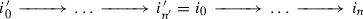of length $$n' + n$$. Then for any pair of composable maps $$g_1$$, $$g_2$$ in $$\Delta I$$, we have a natural map $$c(g_1,g_2):i(g_1)_\cdot * i(g_2)_\cdot \rightarrow i(g_1 \circ g_2)_\cdot$$, and the map (4.2) for the prefibration $$\widetilde{F}$$ is the map $$\alpha (c(g_1,g_2)):g_2^* \circ g_1^* \rightarrow (g_1 \circ g_2)^*$$.

For the reflected prefibration $$\widetilde{F}^\flat$$, the formulas are essentially the same, but we have to count from the other end of a diagram. The fiber $$\widetilde{F}^\flat _{\langle [n],i_\cdot \rangle }$$ is now given by $$\widetilde{F}^\flat _{\langle [n],i_\cdot \rangle } = F(i_n)$$, and the transition functor $$g^*$$ is given by $$g^* = F(i^\flat (g)_\cdot )$$, where $$i^\flat (g)_\cdot$$ is the terminal segment $$i_{g(n')} \rightarrow \dots \rightarrow i_n$$ of the diagram $$i_\cdot$$, of length $$n - g(n')$$. The map (4.2) is again given by $$\alpha (c^\flat (g_1,g_2))$$, where $$c^\flat (g_1,g_2):i^\flat (g_2)_\cdot * i^\flat (g_1)_\cdot \rightarrow i^\flat (g_1 \circ g_2)$$ is the natural map.

Another way to package the same data is by introducing the path 2-category PI of the category I. Its objects are objects $$i \in I$$, 1-morphisms from i to $$i'$$ are pairs $$\langle [n],i_\cdot \rangle$$ with $$i_0=i$$ and $$i'=i_n$$ understood as paths from i to $$i'$$, with composition given by concatenation, and 2-morphisms are maps $$g:\langle [n'],i'_\cdot \rangle \rightarrow \langle [n],i_\cdot \rangle$$ such that $$g(0)=0$$ and $$g(n')=n$$. Then a lax functor F defines an honest 2-functor $$PI \rightarrow {\text {Cat}}$$, $$i \mapsto F(i)$$, $$i_\cdot \mapsto F(i_\cdot )$$, $$g \mapsto \alpha (g)$$, and sending $$\langle [n],i_\cdot \rangle$$ to $$i_0$$ resp. $$i_1$$ and g to $$i(g)_\cdot$$ resp. $$i^\flat (g)_\cdot$$ gives colax functors from $$\Delta I$$ resp. $$(\Delta I)^o$$ to PI.

A special section $$E \in {\text {Sec}}_+(\Delta I,\widetilde{F})$$ is given by a collection of objects $$E(i) = E(\langle ,i \rangle ) \in F(i)$$, $$i \in I$$, and morphisms

\begin{aligned} E(i_\cdot ):E(i_n) \rightarrow F(i_\cdot )(E(i_0)) \end{aligned}
(6.27)

for any $$\langle [n],i_\cdot \rangle \in \Delta I$$, subject to compatibility conditions. In terms of (4.6), we have $$E(i_\cdot ) = E(t)$$, where $$t:\langle ,i_n \rangle \rightarrow \langle [n],i_\cdot \rangle$$ is the natural embedding sending 0 to n. The reflection $$E^\flat$$ is then given by the same objects $$E^\flat (i) = E(i)$$, and the morphisms (6.27) now correspond to the maps (4.6) for the base special maps $$s:\langle ,i \rangle \rightarrow \langle [n],i_\cdot \rangle$$.

Finally, assume given two special sections $$A,B \in {\text {Sec}}_+(\Delta I,\widetilde{F})$$, and let us describe the functor $${\mathcal {H}{} { om}}(A^\flat ,B):\Delta I \rightarrow {\text {Sets}}$$ of Lemma 5.13. For any $$\langle [n],i_\cdot \rangle \in \Delta I$$, we have

\begin{aligned} {\mathcal {H}{} { om}}(A^\flat ,B)(\langle [n],i_\cdot \rangle ) = {\text {Hom}}(A(i_n),F(i_\cdot )(B(i_0))). \end{aligned}
(6.28)

For any map $$g:\langle [n'],i'_\cdot \rangle \rightarrow \langle [n],i_\cdot \rangle$$, let $$\overline{i}(g)_\cdot \subset i_\cdot$$ be the segment of the diagram $$i_\cdot$$ between $$i_{g(0)}$$ and $$i_{g(n')}$$, and let $$\overline{g}:\langle [n'],i'_\cdot \rangle \rightarrow \langle [g(n')-g(0)],\overline{i}(g)_\cdot \rangle$$ be the induced map. Then we have the composition map

\begin{aligned} B(i(g)_\cdot ) \circ A(i^\flat (g)_\cdot ) \circ \alpha (\overline{g}): {\mathcal {H}{} { om}}(A^\flat ,B)(\langle [n'],i'_\cdot \rangle ) \rightarrow {\mathcal {H}{} { om}}(A^\flat ,B)(\langle [n],i_\cdot \rangle ), \end{aligned}

and these maps together with (6.28) give the functor $${\mathcal {H}{} { om}}(A^\flat ,B)$$.

## Reedy categories I

### Ordered categories

Producing model structures on categories of sections of Grothendieck prefibrations is usually a highly non-trivial exercise. This is true even for trivial prefibrations: if we are given a relative category $$\langle \mathcal {C},W \rangle$$ and a small category I, then in general, there is no known construction that would produce a model structure on $$\langle \mathcal {C}^I,W^I \rangle$$ starting from a model structure on $$\langle \mathcal {C},W \rangle$$. However, there are constructions that work either for a restricted class of model structures, or for a restricted class of small categories. Let us recall the latter. We start with a simple special case.

### Definition 7.1

1. (i)

A good filtration on a small category I is a collection of full subcategories $$I_{\le n} \subset I$$, one for any non-negative integer n, such that $$I_{\le n} \subset I_{\le n+1}$$ and $$I = \bigcup I_{\le n}$$.

2. (ii)

A small category I with a good filtration is ordered if

1. (a)

for any integer $$n \ge 0$$, the full subcategory $$I_n \subset I$$ spanned by objects in $$I_{\le n}$$ but not in $$I_{\le n-1}$$ is discrete (that is, all maps are identity maps),

2. (b)

for any morphism $$f:i \rightarrow i'$$, $$i \in I_{\le n}$$ implies $$i' \in I_{\le n}$$, and

3. (c)

for any object $$i \in I$$, the category I(i) of objects $$i' \in I$$ equipped with a map $$f:i \rightarrow i'$$ is finite.

An equivalent way of giving a good filtration is to give a “degree function” $$\deg$$ that associates a non-negative integer n to any object $$i \in I$$, so that $$I_{\le n}$$ is the full subcategory spanned by objects i of degree $$\deg (i) \le n$$.

### Example 7.2

Let V(X) be the partially ordered set of finite subsets in a set X, considered as a small category in the usual way. Then the opposite category $$V(X)^o$$ is ordered, with the degree function given by cardinality.

### Lemma 7.3

Assume given a finite ordered category I and a model category $$\mathcal {C}$$. Then $$\mathcal {C}^I$$ has an injective model structure in the sense of Definition 2.7.

### Sketch of a proof

This is a very well-known fact, but since we will need to generalize it, let us give a sketch of a proof. For any $$n \ge 0$$, an object $$E \in \mathcal {C}^I$$ gives by restriction objects $$E_n \in \mathcal {C}^{I_n}$$, $$E_{\le n} \in \mathcal {C}^{I_{\le n}}$$. The restriction functor $$U:\mathcal {C}^{I_{\le n}} \rightarrow \mathcal {C}^{I_{\le n-1}}$$ has a left-adjoint $$U_\dagger$$ that extends a functor $$E:I_{\le n-1} \rightarrow \mathcal {C}$$ by setting $$E(i)=0$$ for any $$i \in I_n$$, where 0 stands for the initial object. Since $$U \circ U_\dagger \cong \mathsf{Id}$$, $$U_\dagger$$ is fully faithful. Moreover, U also has a right-adjoint $$U^\dagger$$. Indeed, since $$U_\dagger \circ U \cong \mathsf{Id}$$, we have $$U^\dagger \circ U \cong \mathsf{Id}$$, and for any object $$i \in I_n$$, we can set

\begin{aligned} U^\dagger (E)(i) = \mathsf{lim}_{f \in \overline{I(i)}} E(i'), \end{aligned}
(7.1)

where $$\overline{I(i)} \subset I(i)$$ is the full subcategory spanned by non-identical maps $$f:i \rightarrow i'$$. The conditions (a) and (b) of Definition 7.1 (ii) insure that the target $$i'$$ of any such map f lies in $$I_{\le n-1}$$, so that $$E(i')$$ is well-defined, and (c) insures that the category $$\overline{I}(i)$$ is finite, so that the limit exists. Then composing $$U^\dagger$$ with the restriction to $$I_n \subset I_{\le n}$$, we obtain a functor

\begin{aligned} M:\mathcal {C}^{I_{\le n-1}} \rightarrow \mathcal {C}^{I_n}, \end{aligned}

and $$\mathcal {C}^{I_{\le n}}$$ is naturally equivalent to the right comma-category $$\mathsf{R}(M)$$. Observe that by Definition 7.1 (ii) (a), $$\mathcal {C}^{I_n}$$ is just a product of some copies of $$\mathcal {C}$$, thus a model category. Now as in Proposition 3.8 (i), say that a map $$f:E \rightarrow E'$$ in $$\mathcal {C}^I$$ is in F if for any $$n \ge 0$$, the natural map

\begin{aligned} E_n \rightarrow E_n' \times _{M\left( E'_{\le n-1}\right) } M(E_{\le n-1}) \end{aligned}

induced by f via the equivalence $$\mathcal {C}^{I_{\le n}} \cong \mathsf{R}(M)$$ is in F. Then as in the proof of Proposition 3.8 (i), Definition 2.6 (ii), (iii), (iv) are easily checked by induction on n.

$$\square$$

### Remark 7.4

Passing to the opposite categories, we also see that in the assumptions of Lemma 7.3, $$\mathcal {C}^{I^o}$$ has a projective model structure in the sense of Definition 2.7.

We also record right away a couple of properties of injective model structures provided by Lemma 7.3.

### Lemma 7.5

Assume given an ordered category I.

1. (i)

For any model category $$\mathcal {C}$$ and object $$i \in I$$, the functor $$\mathcal {C}^I \rightarrow \mathcal {C}$$ given by evaluation at i is right-derivable with respect to the injective model structure on $$\mathcal {C}^I$$.

2. (ii)

For any model categories $$\mathcal {C}_1$$, $$\mathcal {C}_2$$ and a right-derivable functor $$\Phi :\mathcal {C}_1 \rightarrow \mathcal {C}_2$$ that preserves finite limits, the functor $$\mathcal {C}_1^I \rightarrow \mathcal {C}_2^I$$ induced by $$\Phi$$ is right-derivable,

### Proof

Both claims immediately follow from the inductive description of the injective model structure on $$\mathcal {C}^I$$ given in Lemma 7.3 and the corresponding properties of the model structure of Proposition 3.8 (i). $$\square$$

We note that in Lemma 7.5 (i), the evaluation functor is also trivially left-derivable, and that in Lemma 7.5 (ii), it is essential to require that $$\Phi$$ preserves finite limits.

### Reedy categories

Definition 7.1 and Lemma 7.3 have a remarkable generalization. To state it, recall that a factorization system on a category $$\mathcal {C}$$ consists of two classes of maps $$\langle L,M \rangle$$ in $$\mathcal {C}$$ that are closed under compositions and contain all identity maps, such that any morphism f in $$\mathcal {C}$$ factors as $$f = m \circ l$$, $$l \in L$$, $$m \in M$$, and such a factorization is unique up to a unique isomorphism. For example, for any simplicial set X, special and co-special maps in $$\Delta ^o X$$ in the sense of Definition 5.1 form a factorization system; for more details on this notion, see . For any factorization system $$\langle L,M \rangle$$ on a category $$\mathcal {C}$$, we will denote by $$\mathcal {C}_L$$ resp. $$\mathcal {C}_M$$ the categories with the same objects as $$\mathcal {C}$$ and morphisms that are in L resp. M.

### Definition 7.6

A Reedy category is a small category I equipped with a factorization system $$\langle L,M \rangle$$ and a good filtration in the sense of Definition 7.1 (i) such that both $$I_M$$ and $$I^o_L$$ with the induced filtrations are ordered in the sense of Definition 7.1 (ii).

We note that the notion of a Reedy category is self-dual—for any Reedy category I, the opposite category $$I^o$$ is also a Reedy category, with the same good filtration and $$M^o$$ resp. $$L^o$$ as L resp. M. We also note that for any category $$\mathcal {C}$$, we have $$\left( \mathcal {C}^{I_L}\right) ^o \cong \mathcal {C}^{oI^o_L}$$, so that if $$\mathcal {C}$$ is a model category, then Lemma 7.3 provides natural model structures both on $$\mathcal {C}^{I_M}$$ and on $$\mathcal {C}^{I_L}$$.

### Example 7.7

The category $$\Delta$$ is a Reedy category, with the degree function given by $$\deg ([n])=n$$, and L resp. M consisting of injective resp. surjective maps.

### Example 7.8

More generally, consider the category of simplices $$\Delta X$$ of a simplicial set X, with the degree function $$\deg (\langle [n],x \rangle ) = n$$, and a map f in L resp. M if it is in L resp. M after projecting to $$\Delta$$. Then $$\Delta X$$ is a Reedy category. The only non-trivial thing to check is Definition 7.1 (ii)(c) for the category $$(\Delta X)_M$$; this immediately follows from the fact that for any object $$\langle [n],x \rangle \in \Delta$$, a surjective map $$f:[n] \rightarrow [n']$$ in $$\Delta$$ admits at most one lifting to a map $$\langle [n],x \rangle \rightarrow \langle [n'],x' \rangle$$ in $$\Delta X$$.

### Example 7.9

The category $$\Gamma$$ of finite sets $$\{0,\dots ,n\}$$ and all maps between them is not a Reedy category. Indeed, Definition 7.1 (ii) (a) together with the factorization property imply that $${{\text {Aut}}}(i) = \{\mathsf{id}\}$$ for any object $$i \in I$$ in a Reedy category I.

### Theorem 7.10

Assume given a Reedy category I and a relative category $$\langle \mathcal {C},W \rangle$$ equipped with a model structure $$\langle C,F \rangle$$. Denote by $$\lambda :I_L \rightarrow I$$, $$\mu :I_M \rightarrow I$$ the embedding functors, let $$\widetilde{C}$$ be class of maps f in $$\mathcal {C}^I$$ such that $$\lambda ^*(f)$$ is a cofibration with respect to the projective model structure of Definition 2.7, and let $$\widetilde{F}$$ be the class of maps f such that $$\mu ^*(f)$$ is a fibration with respect to the injective model structure of Definition 2.7. Then $$\langle \widetilde{C},\widetilde{F} \rangle$$ is a model structure on $$\langle \mathcal {C}^I,W^I \rangle$$. $$\square$$

In the literature, Theorem 7.10 is usually formulated as a theorem-construction without first stating Lemma 7.3 (which then appears as a special case, since every ordered category is trivially a Reedy category). While the theorem is due to Reedy , Definition 7.6 is not— only treats the category $$\Delta$$ of Example 7.7 and its opposite $$\Delta ^o$$. The observation that exactly the same proof works in a larger generality was made later, and it is hard to trace when; it definitely appears in , a great reference for the subject, with further references to a book in preparation by Dwyer, Hirshhorn and Kan that, sadly, never appeared (although  uses Reedy model structures in a very essential way).

### Example 7.11

Here are two very basic examples of how one uses Theorem 7.10, both taken out of . Firstly, $$ = \{0,1\}$$ is a Reedy category in two different ways: one can either set $$\deg (0)=0$$, $$\deg (1)=1$$, or the other way around, $$\deg (0)=1$$ and $$\deg (1)=0$$. The two model structures one obtains are the injective and the projective model structure of Definition 2.7. Secondly, for the category $$\mathsf{V}$$ of Sect. 2.2, there are four options: $$\deg (0)=0$$, $$\deg (1)=\deg (2)=1$$, or $$\deg (0)=1$$, $$\deg (1)=\deg (2)=0$$, or $$\deg (1)=2$$, $$\deg (0)=1$$, $$\deg (2)=0$$, or finally $$\deg (1)=0$$, $$\deg (0)=1$$, $$\deg (2)=2$$. The first two options again give the injective and projective model structures, and these give rise to the description of homotopy cartesian and homotopy cocartesian squares in terms of fiber and cofiber squares of Definition 2.8. The other two model structures on $$\mathcal {C}^V$$ are new. Using these structures in the Quillen Adjunction Theorem, one can prove, for example, that a cartesian square (2.4) with fibrant $$Y'$$ is homotopy cartesian as soon as either $$f'$$ or $$g'$$ is a fibration, and dually for cocartesian squares.

The full proof of Theorem 7.10 can be found e.g. in [26, Subsection 5.2], but let us describe the main technical point. One first proves that for any $$n \ge 0$$, any Reedy category I, and any category $$\mathcal {C}$$ with finite limits and colimits, the restriction functor $$U:\mathcal {C}^{I_{\le n}} \rightarrow \mathcal {C}^{I_{\le n-1}}$$ admits both a left and a right-adjoint

\begin{aligned} U_\dagger ,U^\dagger :\mathcal {C}^{I_{\le n-1}} \rightarrow \mathcal {C}^{I_{\le n}}. \end{aligned}

For $$U^\dagger$$, one first restricts to $$I_M$$, defines $$U^\dagger$$ by (7.1), and then observes that for any $$E \in \mathcal {C}^{I_{\le n-1}}$$, $$U^\dagger (E)$$ uniquely extends to $$I_{\le n}$$. For $$U_\dagger$$, one does the same procedure with the opposite categories, restricting to $$I_L$$ instead of $$I_M$$. Explicitly, we have

\begin{aligned} U_\dagger (E)(i) = \mathsf{colim}_{l \in L(i)}E(i'), \quad U^\dagger (i) = \mathsf{lim}_{m \in M(i)}E(i') \end{aligned}
(7.2)

for any $$i \in I_n$$, $$E \in \mathcal {C}^{I_{\le n-1}}$$, where $$L(i) = \overline{I^o_L(i)}^o$$ and $$M(i) = \overline{I_M(i)}$$ are known as the latching and the matching categories of the object i. Both $$U_\dagger$$ and $$U^\dagger$$ are fully faithful, so by adjuntion, we have a map $$U_\dagger \rightarrow U^\dagger$$, and for any $$E \in \mathcal {C}^{I_{\le n}}$$, this maps factors as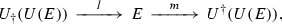where l and m are the adjunction maps. One then observes that the discrete categories $$(I_L)_n$$ and $$(I_M)_n$$ coincide, denotes $$(I_L)_n=(I_M)_n$$ by $$\overline{I}_n \subset I$$, and composes $$U_\dagger$$ and $$U^\dagger$$ with the restriction functor $$R:\mathcal {C}^{I_{\le n}} \rightarrow \mathcal {C}^{\overline{I}_n}$$. This gives two functors

\begin{aligned} L_n = R \circ U_\dagger ,M_n = R \circ U^\dagger :\mathcal {C}^{I_{\le n-1}} \rightarrow \mathcal {C}^{\overline{I}_n}, \end{aligned}

known as the latching and matching functors, and a natural map

\begin{aligned} q:L_n \rightarrow M_n. \end{aligned}
(7.3)

Moreover, for any $$E \in \mathcal {C}^{I_{\le q}}$$, the quadruple $$\langle U(E),R(E),R(l),R(m) \rangle$$ is an object of the two-sided comma-category $$\mathsf{G}(L_n,M_n,q)$$ of Definition 3.10, so that we have a natural functor

\begin{aligned} \mathcal {C}^{I_{\le n}} \rightarrow \mathsf{G}(L_n,M_n,q). \end{aligned}
(7.4)

Then one proves the following crucial fact: the functor (7.3) is an equivalence of categories.

With this fact, the proof of Theorem 7.10 proceeds by induction on the degree, as in Lemma 7.3. In our context, one can easily deduce from (7.2) that the latching functors $$L_n$$ resp. $$M_n$$ are left resp. right-derivable, and then it suffices to apply Proposition 3.12 (i). The usual proofs spell things out more explicitly (and in fact, our proof of Proposition 3.12 is extracted from the standard proofs of Theorem 7.10).

### Balzin theorem

A very useful result discovered recently by Balzin  generalizes Theorem 7.10 to non-trivial families of model categories.

### Definition 7.12

A prefibration $$\pi :\mathcal {C}\rightarrow I$$ over a Reedy category $$\langle I,L,M \rangle$$ is good if

1. (i)

for any morphism $$l:i' \rightarrow i$$ in L, the transition functor $$l^*:\mathcal {C}_i \rightarrow \mathcal {C}_{i'}$$ has a left-adjoint $$l_!:\mathcal {C}_{i'} \rightarrow \mathcal {C}_i$$, and

2. (ii)

if we are given another morphism $$f:i \rightarrow i''$$ in I, then the natural map $$l^* \circ f^* \rightarrow (f \circ l)^*$$ is an isomorphism.

### Remark 7.13

If I is an ordered category, so that L consists of identity maps, then every prefibration $$\mathcal {C}\rightarrow I$$ is good.

### Remark 7.14

If $$\mathcal {C}\rightarrow I$$ is a Grothendieck fibration, then the second condition of Definition 7.12 is automatic.

### Definition 7.15

A model prefibration $$\mathcal {C}\rightarrow I$$ over a category I is a prefibration equipped with classes of fiberwise maps W, C, F such that

1. (i)

for any object $$i \in I$$, C, F and W turn the fiber $$\mathcal {C}_i$$ into a model category, and

2. (ii)

for any morphism $$f:i' \rightarrow i$$ in I, the transition functor $$f^*:\mathcal {C}_i \rightarrow \mathcal {C}_{i'}$$ is right-derivable in the sense of Definition 3.1.

### Definition 7.16

A functor $$\gamma :I' \rightarrow I$$ between Reedy categories $$\langle I,L,M \rangle$$, $$\langle I',L',M'\rangle$$ is a Reedy functor if it sends $$L'$$ to L and $$M'$$ to M.

For any Reedy functor $$\gamma :I' \rightarrow I$$ and a prefibration $$\mathcal {C}$$ over I, $$\gamma ^*\mathcal {C}$$ is good if $$\mathcal {C}$$ is good, and carries a natural structure of a model prefibration if so does $$\mathcal {C}$$. In particular, this applies to the natural embeddings

\begin{aligned} \lambda :I_L \rightarrow I, \qquad \mu :I_M \rightarrow I, \end{aligned}
(7.5)

since both are tautologically Reedy functors.

### Theorem 7.17

Assume given a good model prefibration $$\mathcal {C}\rightarrow I$$ over a Reedy category I. Moreover, assume that either of the following two conditions holds:

1. (i)

the restriction $$\mu ^*\mathcal {C}\rightarrow I_M$$ is a Grothendieck fibration whose transition functors preserves finite limits,

2. (ii)

for any object $$i \in I$$, the matching category M(i) has an initial object.

Then $${\text {Sec}}(I,\mathcal {C})$$ with pointwise weak equivalences has a natural model structure. If a map f in $${\text {Sec}}(I,\mathcal {C})$$ is a cofibration or a fibration, then so is f(i) for any $$i \in I$$, so that the evaluation functor (4.7) is left and right-derivable. If I is discrete, then conversely, a map f is a cofibration or fibration if so is f(i) for any object $$i \in I$$. In general, f is a cofibration iff $$\lambda ^*(f)$$ is a cofibration, and a fibration iff $$\mu ^*(f)$$ is a fibration.

We note for any Reedy category I, $$(I_M)_L=(I_L)_M$$ is discrete, so that as in Theorem 7.10, the model structure with the properties listed in Theorem 7.17 is unique. For any fixed model category $$\mathcal {C}$$ and Reedy category I, $$\mathcal {C}\times I \rightarrow I$$ is trivially a Reedy model prefibration, and $${\text {Sec}}(I,\mathcal {C}\times I) \cong \mathcal {C}^I$$, so that Theorem 7.17 generalizes Theorem 7.10. The simplest new case of Theorem 7.17 appears for $$I=$$, and this is exactly the case that we have considered in Proposition 3.8.

To prove Theorem 7.17, Balzin generalizes the inductive description of $$\mathcal {C}^I$$ given in Sect. 7.2. Namely, for any integer n, denote by $$\tau _{\le n}:I_{\le n} \rightarrow I$$, $$\overline{\tau }_n:\overline{I}_n \rightarrow I$$ the embedding functors, and for any good prefibration $$\mathcal {C}\rightarrow I$$, consider the restriction functor $$U:{\text {Sec}}(I_{\le n},\tau _{\le n}^*\mathcal {C}) \rightarrow {\text {Sec}}(I_{\le n-1},\tau _{\le n-1}^*\mathcal {C})$$. Then Balzin proves the following generalization of the equivalence (7.4).

### Lemma 7.18

\begin{aligned} U_\dagger ,U^\dagger :{\text {Sec}}\left( I_{\le n-1},\tau _{\le n-1}^*\mathcal {C}\right) \rightarrow {\text {Sec}}\left( I_{\le n},\tau _{\le n}^*\mathcal {C}\right) . \end{aligned}

Moreover, denote $$L_n = \overline{\tau }_n^* \circ U_\dagger$$, $$M_n = \overline{\tau }_n^* \circ U^\dagger$$, and let $$q:L_n \rightarrow M_n$$ be the map induced by the natural map $$U_\dagger \rightarrow U^\dagger$$. Then we have an equivalence of categories

\begin{aligned} {\text {Sec}}\left( I_{\le n},\tau _{\le n}^*\mathcal {C}\right) \cong \mathsf{G}(L_n,M_n,q). \end{aligned}
(7.6)

Explicitly, the adjoint functors in Lemma 7.18 are given by modifying (7.2) to

\begin{aligned} U_\dagger (E)(i) = \mathsf{colim}_{l \in L(i)}l_!E(i'), \quad U^\dagger (i) = \mathsf{lim}_{m \in M(i)}m^*E(i'), \end{aligned}
(7.7)

where $$l_!$$ is the adjoint functor of Definition 7.12 (i). In particular, we see that we have natural isomorphisms

\begin{aligned} \mu ^* \circ U^\dagger \cong U^\dagger \circ \mu ^*, \qquad \lambda ^* \circ U_\dagger \cong U_\dagger \circ \lambda ^*, \end{aligned}
(7.8)

where $$U_\dagger$$ resp. $$U^\dagger$$ in the right-hand side are the corresponding adjoint functors for the categories $$I_L$$ resp. $$I_M$$. Moreover, if we have another good model prefibration $$\mathcal {C}' \rightarrow I$$ and functor $$\Phi :\mathcal {C}\rightarrow \mathcal {C}'$$ cartesian over I and such that $$\Phi (i)$$, $$i \in I$$ preserve finite limits, then we have a natural isomorphism

\begin{aligned} \Phi ' \circ U^\dagger \cong U^\dagger \circ \Phi . \end{aligned}
(7.9)

One can deduce Theorem 7.17 from Lemma 7.18 by applying Proposition 3.12, but there is a hitch: one has to insure that when $$\mathcal {C}$$ is a model prefibration, the latching and matching functors $$L_n$$, $$M_n$$ are left resp. right-derivable. In the standard Reedy case, this follows from (7.2). Indeed, for any $$i \in I$$, the matching category M(i) is ordered, every fibration in $${\text {Sec}}(I_{\le n-1},\tau _{\le n-1}^*\mathcal {C})$$ restricts to a fibration in $$\mathcal {C}_i^{M(i)}$$ with respect to the injective model structure, and $$\mathsf{lim}_{M(i)}$$ is a right-Quillen functor, thus sends fibrations to fibrations. The argument for $$L_n$$ is dual.

In the case (i) of Theorem 7.17, the same argument works, and since the functors $$l_!$$ preserve colimits by adjunction, it also works for $$L_n$$ in the general case. For $$M_n$$, there is a problem: a fibration in $${\text {Sec}}(I_{\le n-1},\mathcal {C})$$ in general only restricts to a pointwise fibration in $$\mathcal {C}_i^{M(i)}$$ and not necessarily to a fibration with respect to the injective model structure. However, under the assumption (ii) of Theorem 7.17, taking $$\mathsf{lim}_{M(i)}$$ amounts to evaluating at the initial object of the category M(i), so that pointwise fibrations are good enough.

### Remark 7.19

In fact, what Balzin proves in  is even more general than Theorem 7.17: instead of our notion of a good prefibration, he introduces a more general notion of a semifibration over a category with a factorization system. This treats both sides of the factorization system on an equal footing, and in the Reedy case, contains exactly enough structure to define the functors (7.7) and the map (7.3). We will not need this larger generality.

### Matching expansions

We note that case (i) of Theorem 7.17 is only very slightly stronger than known results of Simpson and Hirschowitz . The real novelty is in the case (ii), and at a first glance, the condition imposed on the Reedy category I is extremely restrictive. However, it turns out that it can always be satisfied by enlarging I.

Assume given a Reedy category $$\langle I,L,M,\deg \rangle$$, and consider the simplicial expansion $$\Delta ^M_L I$$ of Definition 5.5. Recall that explicitly, objects in $$\Delta ^M_L I$$ are pairs $$\langle [n],i_\cdot \rangle$$ of an object $$[n] \in \Delta$$ and a diagram (5.3) in $$I_M$$, so that for any $$0 \le j < j' \le n$$ we have a natural map $$i_j \rightarrow i_{j'}$$. Since the class M is closed under compositions, the forgetful functor $$\Delta ^M_L I \rightarrow \Delta$$ is a Grothendieck fibration. Let $$\pi :(\Delta ^M_L I)^\perp \rightarrow \Delta ^o$$ be the transpose Grothendieck cofibration. Recall that by definition, $$(\Delta ^L_M I)^\perp$$ has the same objects $$\langle [n],i_\cdot \rangle$$ as $$\Delta ^L_M I$$, and for any morphism f in $$\Delta ^o$$, the transition functor $$f_!$$ is canonically identified with the transition functor $$(f^o)^*$$, where $$f^o$$ is f considered as a morphism in $$\Delta$$. A morphism from $$\langle [n'],i'_\cdot \rangle$$ to $$\langle [n],i_\cdot \rangle$$ in $$(\Delta ^L_M I)^\perp$$ is given by a map $$f:[n] \rightarrow [n']$$ in $$\Delta$$ and a collection of maps $$l_j:i'_{f(j)} \rightarrow i_j$$, $$0 \le j \le n$$ in the class L such that the diagram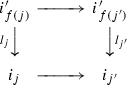commutes for every $$0 \le j \le j' \le n$$. Sending $$\langle [n],i_\cdot \rangle$$ to $$i_0$$ gives a functor

\begin{aligned} \left( \Delta ^L_M\right) ^\perp \rightarrow I. \end{aligned}
(7.10)

Say that an object $$\langle [n],i_\cdot \rangle \in (\Delta ^L_M I)^\perp$$ is non-degenerate if all the maps $$i_j \rightarrow i_{j'}$$, $$j < j'$$ are not identity maps (equivalently, $$\deg i_0> \dots > \deg i_n$$).

### Definition 7.20

The matching expansion M(I) of the Reedy category I is the full subcategory $$M(I) \subset (\Delta ^M_L I)^\perp$$ spanned by non-degenerate objects.

Note that every diagram (5.3) can be reduced to a non-degenerate one by contracting all the identity maps $$i_j \rightarrow i_j$$ that occur. In terms of the Grothendieck fibration $$\Delta ^L_M I \rightarrow \Delta$$, this means that for any $$\langle [n],i_\cdot \rangle$$, there exists a unique surjective map $$[n] \rightarrow [n']$$ and a non-degenerate object $$\langle [n'],i'_\cdot \rangle$$ such that $$f^*i'_\cdot = i_\cdot$$. We call $$\langle [n'],i'_\cdot \rangle$$ the normalization of $$\langle [n],i_\cdot \rangle$$. Sending an object to its normalization then gives a functor

\begin{aligned} N:\left( \Delta ^L_M I\right) ^\perp \rightarrow M(I) \end{aligned}
(7.11)

right-adjoint to the embedding $$M(I) \subset (\Delta ^L_M)^\perp$$. We can also restrict the Grothendieck cofibration $$\pi$$ and the functor (7.10) to $$M(I) \subset (\Delta ^L_M)^\perp$$ and obtain functors

\begin{aligned} \pi :M(I) \rightarrow \Delta ^o, \qquad \rho :M(I) \rightarrow I. \end{aligned}
(7.12)

Since the normalization of a diagram (5.3) has the same initial object $$i_0$$, we have $$\rho \circ N \cong \rho$$.

### Lemma 7.21

The functor $$\rho$$ of (7.12) is a prefibration.

### Proof

Assume given an object $$\langle [n],i_\cdot \rangle$$ in M(I) and a map $$g:i_0' \rightarrow i_0 = \rho (\langle [n],i_\cdot \rangle )$$ in the category I. Then for any j, $$0 \le j \le n$$, we can compose g with the natural map $$i_0 \rightarrow i_j$$ and obtain a map $$g_j:i_0' \rightarrow i_j$$. Factorize these maps as $$g_j = l_j \circ m_j$$, with $$m_j:i_0' \rightarrow i'_{j+1}$$ in M and $$l_j:i'_{j+1} \rightarrow i_j$$ in L, and consider the induced diagram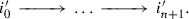Since L and M form a factorization system on I, all the maps in this diagram are in M, so that it gives an object $$\langle [n+1],i'_\cdot \rangle$$ in $$(\Delta ^L_M I)^\perp$$. Moreover, if we let $$f:[n] \rightarrow [n+1]$$ be the shift map $$j \mapsto j+1$$, then f with the morphisms $$l_j$$ defines a map

\begin{aligned} \widetilde{g}:\langle [n+1],i'_\cdot \rangle \rightarrow \langle [n],i_\cdot \rangle \end{aligned}

in the category $$(\Delta ^L_M I)^\perp$$. We have $$\rho (\widetilde{g})=g$$, and $$\widetilde{g}$$ is obviously cartesian with respect to the projection (7.10). If $$i'_\cdot$$ is non-degenerate, then $$\widetilde{g}$$ gives the desired cartesian lifting of the map g. If not, replace it with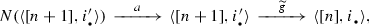where N is the normalization functor (7.11), and a is the adjunction map. Definition 7.12 (ii) is then immediately obvious. $$\square$$

We note that it is easy to describe the fibers $$M(I)_i$$, $$i \in I$$ of the prefibration $$\rho$$ of Lemma 7.21. By definition, the fiber $$M(I)_i \subset M(I)$$ is the full subcategory spanned by $$\langle [n],i_\cdot \rangle$$ with $$i_0=i$$. Consider the matching category M(i) of the object $$i \in I$$ with its simplicial replacement $$\Delta M(i)$$, and let $$\overline{\Delta } M(i) \subset \Delta M(i)$$ be the full subcategory spanned by non-degenerate simplices. Then we have a natural identification

\begin{aligned} M(I)_i^o \cong \left( \overline{\Delta } M(i)\right) ^<. \end{aligned}
(7.13)

The initial object o that we add to $$\overline{\Delta } M(i)$$ to form $$\left( \overline{\Delta } M(i)\right) ^<$$ corresponds to the terminal object $$\langle ,i \rangle \in M(I)_i$$. Note that since M(i) is a finite category, $$\overline{\Delta } M(i)$$ is also finite, and then so is the fiber $$M(I)_i$$.

Now define the degree function on M(I) by

\begin{aligned} \deg (i) = 2\deg (\rho (i))^2 - \deg (\pi (i)), \qquad i \in M(I), \end{aligned}
(7.14)

and define classes of morphisms L, M in M(I) by

1. (i)

$$f \in L$$ iff $$\pi (f)^o$$ is a special map in $$\Delta$$ in the sense of Definition 5.1,

2. (ii)

$$f \in M$$ iff $$\pi (f)^o$$ is co-special and f is cocartesian with respect to $$\pi$$.

### Example 7.22

Let $$I=$$, with the degree function $$\deg (0)=1$$, $$\deg (1)=0$$. Then $$M(I) \cong \mathsf{V}$$, with the degree function $$\deg (1)=0$$, $$\deg (0)=1$$, $$\deg (2)=2$$ (as in Example 7.11).

### Proposition 7.23

The matching expansion M(I) of a Reedy category I with the classes L, M and the degree function as above is a Reedy category, and it satisfies the condition (ii) of Theorem 7.17.

### Proof

Since $$\pi :(\Delta ^L_M I)^\perp \rightarrow \Delta ^o$$ is a Grothendieck cofibration, and special and co-special maps form a factorization system in $$\Delta$$, any map $$f:c \rightarrow c'$$ in $$(\Delta ^L_M I)^\perp$$ factors as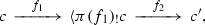(7.15)

where $$\pi (f_1)^o$$ resp. $$\pi (f_2)^o$$ is cospecial resp. special, and $$f_1$$ is cocartesian over $$\Delta ^o$$. Such a factorization is unique up to a unique isomorphism. For any injective map $$g:[n] \rightarrow [n']$$ in $$\Delta$$, the transition functor $$g^*$$ of the Grothendieck fibration $$\Delta ^L_M I \rightarrow \Delta$$ preserves the non-degeneracy condition. Since co-special maps are injective, this applies to $$(\pi (f_1)^o)^* \cong \pi (f_1)_!$$. Therefore if $$c,c' \in M(I)$$, then $$\pi (f_1)_!c \in M(I)$$, so that $$f_1 \in M$$, $$f_2 \in L$$, and L, M do form a factorization system on M(I).

Next, observe that since the degree function on I is by definition non-negative, we have $$\deg (i_0) \ge n$$ for any non-degenerate diagram (5.3), so that the degree function (7.14) is also non-negative. Moreover, the degree function (7.14) is chosen in such a way that the induced order on objects $$c \in M(I)$$ is lexicographic: $$\deg (c) < \deg (c')$$ iff either $$\deg (\rho (c)) < \deg (\rho (c'))$$, or $$\deg (\rho (c)) = \deg (\rho (c'))$$ and $$\deg (\pi (c')) < \deg (\pi (c))$$.

For any object $$c = \langle [n],i_\cdot \rangle \in M(I)$$, the matching category M(c) by definition consists of cocartesian liftings $$c \rightarrow f^o_!c$$ of the maps $$f^o$$ opposite to non-identity cospecial maps $$f:[n'] \rightarrow [n]$$. Thus it is equivalent to the category $$[n-1]$$. In particular, it is finite and satisfies (ii) of Theorem 7.17. Moreover, for any such map $$f:[n'] \rightarrow [n]$$, we have $$\deg (\rho (f^o_!c)) = \deg (i_{f(0)}) < \deg (i_0) = \deg (\rho (c))$$, so that the cocartesian lifting of $$f^o$$ lowers the degree.

For the latching category, observe that in fact a map $$f:c \rightarrow c'$$ in M(I) is in the class L if and only if $$\rho (f)$$ is the class L. Then if $$\rho (f)$$ is not an identity map, we have $$\deg (\rho (c)) < \deg (\rho (c'))$$, and if $$\rho (f)$$ is an identity map but f is not, then $$\deg (\rho (c))=\deg (\rho (c'))$$ but $$\deg (\pi (c)) > \deg (\pi (c'))$$, so that in any case $$\deg (c) < \deg (c')$$. Moreover, the latching category L(c) is the total space of the prefibration $$\rho :L(c) \rightarrow L(\rho (c))$$ with fibers (7.13), the fibers are finite, and $$L(\rho (c))$$ is also finite by assumption. Therefore L(c) is finite, and this finishes the proof. $$\square$$

### Derived sections

As a corollary of Proposition 7.23, we see that for any good model prefibration $$\mathcal {C}$$ over a Reedy category I, the category $${\text {Sec}}(M(I),\rho ^*\mathcal {C})$$ has a natural model structure provided by Theorem 7.17. We have the pullback functor

\begin{aligned} \rho ^*:{\text {Sec}}(I,\mathcal {C}) \rightarrow {\text {Sec}}(M(I),\rho ^*\mathcal {C}), \end{aligned}
(7.16)

and for any $$i \in I$$, it restricts to the tautological functor

\begin{aligned} \rho ^*(i):\mathcal {C}_i \rightarrow \mathcal {C}_i^{M(I)_i}. \end{aligned}
(7.17)

The fibers $$M(I)_i$$ of the prefibration $$\rho$$ are given by (7.13), and in particular, they have terminal objects. Evaluating at this terminal object gives a left-adjoint $$\rho _!(i)$$ to the functor (7.17), and we obviously have $$\rho _!(i) \circ \rho ^*(i) \cong \mathsf{Id}$$, so that $$\rho ^*(i)$$ is fully faithful. Moreover, both $$\rho ^*(i)$$ and $$\rho _!(i)$$ preserve weak equivalences, thus descend to an adjoint pair of functors on the homotopy categories, and therefore the functor $${\text {Ho}}(\rho ^*(i))$$ is fully faithful as well.

One can ask whether the same holds globally over I. More precisely, let us introduce the following.

### Definition 7.24

A section $$\sigma \in {\text {Sec}}(I,\mathcal {C})$$ of a model prefibration $$\mathcal {C}$$ over a small category $$\mathcal {C}$$ is homotopy cartesian along a map f if the corresponding map (4.6) is a weak equivalence.

### Definition 7.25

For any good model prefibration $$\mathcal {C}$$ over a Reedy category I with the matching expansion $$\rho :M(I) \rightarrow I$$, a derived section $$\sigma$$ of $$\mathcal {C}$$ over I is a section $$\sigma \in {\text {Sec}}(M(I),\rho ^*\mathcal {C})$$ that is homotopy cartesian along all maps f in M(I) vertical with respect to $$\rho$$. The full subcategory in $${\text {Sec}}(M(I),\rho ^*\mathcal {C})$$ spanned by derived sections is denoted by $${\text {Sec}}_{\rho }(M(I),\rho ^*\mathcal {C})$$.

Then the embedding $${\text {Sec}}_{\rho }(M(I),\rho ^*\mathcal {C}) \rightarrow {\text {Sec}}(M(I),\rho ^*\mathcal {C})$$ is obviously an example of the model embedding in the sense of Definition 2.10. We denote by

\begin{aligned} {\text {DSec}}(I,\mathcal {C}) = {\text {Ho}}({\text {Sec}}_{\rho }(M(I),\rho ^*\mathcal {C})) \end{aligned}
(7.18)

the homotopy category of derived sections, and we can ask whether the pullback functor

\begin{aligned} {\text {Ho}}({\text {Sec}}(I,\mathcal {C})) \rightarrow {\text {DSec}}(I,\mathcal {C}) \end{aligned}
(7.19)

is an equivalence of categories—so that, lacking a model structure on $${\text {Sec}}(I,\mathcal {C})$$, we at least have a model embedding.

Unfortunately, we do not know whether this statement is always true. So, for a general good model prefibration $$\mathcal {C}/I$$, we simply take $${\text {DSec}}(I,\mathcal {C})$$ as a correct replacement for $${\text {Ho}}({\text {Sec}}(I,\mathcal {C}))$$. As a justification for this, let us prove that the two agree in the cases covered by Theorem 7.17,

### Proposition 7.26

Assume given a good model prefibration $$\mathcal {C}$$ over a Reedy category I that satisfies either of the two conditions of Theorem 7.17. Then the functor (7.19) is an equivalence of categories.

### Proof

In the case (ii) of Theorem 7.17, note that by induction, the matching category M(i) for any $$i \in I$$ is an ordinal category [n]. Then the fiber $$M(I)_i$$ of the prefibration $$\rho$$ has an initial object corresponding to the longest non-degenerate diagram (5.3). Sending i to this initial object defines a section $$\sigma :I \rightarrow M(I)$$ of the prefibration $$\rho$$, and $$\sigma$$ is left-adjoint to $$\rho$$. Therefore $$\sigma ^*$$ is right-adjoint to the fully faithful functor (7.16). Since both functors $$\sigma ^*$$ and $$\rho ^*$$ obviously preserve weak equivalences, they induce an adjoint pair of functors on homotopy categories, and since $$\rho \circ \sigma = \mathsf{id}$$, the functor $${\text {Ho}}(\rho ^*):{\text {Ho}}({\text {Sec}}(I,\mathcal {C})) \rightarrow {\text {Ho}}({\text {Sec}}(M(I),\rho ^*\mathcal {C}))$$ induced by (7.16) is fully faithful. Moreover, for any derived section s of $$\mathcal {C}$$ over I, the adjunction map $$\rho ^*\sigma ^*s \rightarrow s$$ is a pointwise weak equivalence, so that it lies in the image of the fully faithful functor $${\text {Ho}}(\rho ^*)$$.

In the case (i), we no longer have the section $$\sigma$$. However, for any i, since the fiber $$M(I)_i$$ is finite, the functor $$\rho ^*(i)$$ of (7.17) has a right-adjoint $$\rho _*(i) = \mathsf{lim}_{M(I)_i}$$, and since the transition functors of the prefibration $$\mathcal {C}\rightarrow I$$ preserve limits, we are in the situation of Lemma 4.16. Therefore the functors $$\rho _*(i)$$ together define a functor

\begin{aligned} \rho _*:{\text {Sec}}(M(I),\rho ^*\mathcal {C}) \rightarrow {\text {Sec}}(I,\mathcal {C}) \end{aligned}

right-adjoint to $$\rho ^*$$, and for any $$i \in I$$, we have $$\mathsf{ev}_i \circ \rho _* \cong \rho _*(i) \circ \varepsilon (i)^*$$, where $$\mathsf{ev}_i$$ is the evaluation functor (4.7), and $$\varepsilon (i):M(I)_i \rightarrow M(I)$$ is the embedding of the fiber $$M(I)_i$$. Moreover, the adjunction map $$\mathsf{Id}\rightarrow \rho _* \circ \rho ^*$$ is an isomorphism at every $$i \in I$$, thus an isomorphism, so that $$\rho ^*$$ is fully faithful

To see what happens on the level of homotopy categories, it is convenient to change the Reedy structure on the matching expansion M(I), by replacing (7.14) with $$\deg (i) = 2\deg (\rho (i))^2 + \deg (\pi (i))$$, and redefining classes L and M by saying that $$f \in M$$ iff $$\rho (f) \in M$$, and $$f \in L$$ iff $$\rho (f) \in L$$ and f is cartesian with respect to $$\rho$$. Then by essentially the same argument as in Proposition 7.23, this turns M(I) into a Reedy category, and while it no longer satisfies the condition (ii) of Theorem 7.17, the model prefibration $$\rho ^*\mathcal {C}$$ is still good and still satisfies the condition (i). Thus Theorem 7.17 provides a new model structure on $${\text {Sec}}(M(I),\rho ^*\mathcal {C})$$, with the same class of weak equivalences and the same homotopy category. However, now for any $$i \in M(I)$$, the projection $$\rho$$ induces an equivalence of latching categories $$L(i) \cong L(\rho (i))$$, so that the pullback functor $$\rho ^*$$ commutes with latching functors and is therefore left-derivable. Then $$\rho _*$$ is right-derivable by adjunction, and to finish the proof as in the case (ii), it suffices to check that the adjunction map $$\mathsf{Id}\rightarrow R^\cdot \rho _* \circ L^\cdot \rho ^*$$ is an isomorphism. Morever, for every $$i \in I$$, we already know that $$\rho ^*(i) \cong L^\cdot \rho ^*(i)$$ is fully faithful, so it actually suffices to prove that on the derived level, we still have $$\mathsf{ev}_i \circ R^\cdot \rho _* \cong R^\cdot \rho _*(i) \circ \varepsilon (i)^*$$. This amounts to checking that

\begin{aligned} \varepsilon (i)^*:{\text {Sec}}(M(I),\rho ^*\mathcal {C}) \rightarrow \mathcal {C}_i^{M(I)_i} \end{aligned}

is right-derivable with respect to the new model structure on $${\text {Sec}}(M(I),\rho ^*\mathcal {C})$$ and the injective model structure on $$\mathcal {C}_i^{M(I)_i}$$.

To do this, first note that with the new Reedy structure on M(I), we have $$M(I)_M=M(I_M)$$, so by Theorem 7.17, we can replace I with $$I_M$$ and assume right away that I is an ordered category (and $$\mathcal {C}$$ is then a Grothendieck fibration). Moreover, for any discrete Grothendieck cofibration $$\kappa :I' \rightarrow I$$, $$I'$$ is also an ordered category, and $$M(\kappa ):M(I') \rightarrow M(I)$$ is a discrete Grothendieck cofibration that induces equivalences of matching categories. Then $$M(\kappa )^*$$ commutes with matching functors, thus is right-derivable. Therefore we can replace I with the category I(i) and assume that $$i \in I$$ is the initial object. For the final reduction, note that we then have the constant functor $$s:I \rightarrow I$$ with value $$i \in I$$ and the map $$a:s \rightarrow \mathsf{id}$$, and a induces a functor $$a^*:\mathcal {C}\rightarrow \mathcal {C}_i \times I$$ to the constant Grothendieck fibration with fiber $$\mathcal {C}_i$$. Moreover, this functor is cartesian, and for any $$i' \in I$$, $$a(i')$$ commutes with finite limits. Then by (7.9), $$a^*$$ commutes with matching functors, thus is right-derivable, so that we may assume that $$\mathcal {C}\rightarrow I$$ is a constant Grothendieck fibration with fiber $$\mathcal {C}_i$$, and $${\text {Sec}}(M(I),\rho ^*\mathcal {C})$$ is then the functor category $$\mathcal {C}_i^{M(I)}$$ with the injective model structure. But since $$\rho :M(I) \rightarrow I$$ is a prefibration, the embedding $$\varepsilon (i):M(I)_i \rightarrow M(I)$$ of the fiber over the initial object i admits a right-adjoint functor $$\varepsilon (i)^\dagger$$, and the pullback functor $$(\varepsilon (i)^\dagger )^*$$ is then left-adjoint to $$\varepsilon (i)^*$$. Since any pullback functor is trivially left-derivable for injective model structures, $$\varepsilon (i)^*$$ is right-derivable by adjunction. $$\square$$

## Reedy categories II

### Derived sections of stable pairs

In the situation of stable model pairs, it is easy to show that Balzin model structure of Theorem 7.17 also gives a stable model pair (this also works in the classical Reedy case).

### Definition 8.1

A stable model prefibration $$\langle \mathcal {C},\mathcal {C}' \rangle$$ over a category I is a model prefibration $$\mathcal {C}' \rightarrow I$$ equipped with a full subcategory $$\mathcal {C}\subset \mathcal {C}'$$ such that for any $$i \in I$$, $$\langle \mathcal {C}_i,\mathcal {C}'_i \rangle$$ is a stable model pair, and for any morphism $$f:i' \rightarrow i$$ in I, the transition functor $$f^*:\mathcal {C}'_i \rightarrow \mathcal {C}'_{i'}$$ is a stable right-derivable functor between stable model pairs. A stable model prefibration $$\langle \mathcal {C},\mathcal {C}' \rangle$$ over a Reedy category I is good if $$\mathcal {C}' \rightarrow I$$ is good in the sense of Definition 7.12.

For any stable model prefibration $$\langle \mathcal {C},\mathcal {C}' \rangle$$ over I, the homotopy categories $${\text {Ho}}(\mathcal {C}_i)$$ of the fibers $$\mathcal {C}_i$$, $$i \in I$$ together with the functors $${\text {Ho}}(\mathcal {C}_{i'}) \rightarrow {\text {Ho}}(\mathcal {C}_i)$$, $$f:i \rightarrow i'$$ induced by the transition functors of the prefibration $$\mathcal {C}'$$ form a prefibration over I. We will denote it by

\begin{aligned} {\text {Ho}}(\mathcal {C}/I) \rightarrow I. \end{aligned}
(8.1)

If the stable model prefibration $$\langle \mathcal {C},\mathcal {C}' \rangle$$ is good, then the prefibration (8.1) is also good.

### Proposition 8.2

Assume given a good stable model prefibration $$\langle \mathcal {C},\mathcal {C}' \rangle$$ over a Reedy category I with the matching expansion $$\rho :M(I) \rightarrow I$$, and consider the full subcategory

\begin{aligned} {\text {Sec}}_\rho (M(I),\rho ^*\mathcal {C}) = {\text {Sec}}_\rho (M(I),\rho ^*\mathcal {C}') \cap {\text {Sec}}(M(I),\rho ^*\mathcal {C}) \end{aligned}
(8.2)

in $${\text {Sec}}(M(I),\rho ^*\mathcal {C}')$$ spanned by derived sections of Definition 7.25 that take values in $$\rho ^*\mathcal {C}\subset \rho ^*\mathcal {C}'$$. Then $$\langle {\text {Sec}}_\rho (M(I),\rho ^*\mathcal {C}),{\text {Sec}}(M(I),\rho ^*\mathcal {C}') \rangle$$ is a stable model pair with respect to the model structure on $${\text {Sec}}(M(I),\rho ^*\mathcal {C}')$$ provided by Theorem 7.17.

### Proof

Consider first the full subcategory $${\text {Sec}}(M(I),\rho ^*\mathcal {C}) \subset {\text {Sec}}(M(I),\rho ^*\mathcal {C}')$$. Since fibrations, cofibrations, and cartesian and cocartesian squares in the category $${\text {Sec}}(M(I),\mathcal {C}')$$ are defined inductively via Lemma 7.18, to prove that $$\langle {\text {Sec}}(M(I),\rho ^*\mathcal {C}),{\text {Sec}}(M(I),\rho ^*\mathcal {C}') \rangle$$ is a stable model pair, it suffices to prove that for every integer n, the matching and latching functors

\begin{aligned} L_n,M_n:{\text {Sec}}(M(I)_{\le n-1},\tau _{\le n-1}\rho ^*\mathcal {C}') \rightarrow {\text {Sec}}\left( \overline{M(I)}_n,\overline{\tau }_n^*\rho ^*\mathcal {C}'\right) \end{aligned}

of (7.7) are stable, and apply Proposition 3.12 (ii).

For $$L_n$$, this is clear: the functors $$l_!$$ in (7.7) are left-Quillen by Definition 7.12, so that $$L_n$$ is also left-Quillen, and for any $$i \in M(I)$$, $$\mathcal {C}_i \subset \mathcal {C}'_i$$ is closed under homotopy colimits by Definition 2.12 (ii), $$L^\cdot L_n$$ sends sections of $$\rho ^*\mathcal {C}\subset \rho ^*\mathcal {C}'$$ into sections of $$\rho ^*\mathcal {C}$$.

For $$M_n$$, recall that the Reedy category M(I) satisfies the assumption (ii) of Theorem 7.17, so that taking the limit in (7.7) amounts to evaluating at the initial object of the category M(i). Proposition 3.12 immediately implies by induction that homotopy cartesian squares in $${\text {Sec}}(M(I)_{\le n},\tau ^*_{\le n}\rho ^*\mathcal {C}')$$ are pointwise homotopy cartesian, and since the transition functors $$f^*$$ in (7.7) are stable by Definition 8.1, the matching functor $$M_n$$ is also stable.

But now, by virtue of (8.2), $${\text {Sec}}_\rho (M(I),\rho ^*\mathcal {C}) \subset {\text {Sec}}(M(I),\rho ^*\mathcal {C}')$$ satisfies Definition 2.12 (i) automatically, so that it remains to check Definition 2.12 (ii). This is obvious: by Proposition 3.12, a square in the category $${\text {Sec}}(M(I),\rho ^*\mathcal {C})$$ is homotopy cartesian or cocartesian if and only if this holds pointwise, and the transition functor $$f^*$$ for any map f in M(I) vertical with respect to $$\rho$$ is an equivalence of categories, thus sends homotopy cartesian squares to homotopy cartesian squares. $$\square$$

Proposition 8.2 and Proposition 2.20 show that for any good stable model prefibration $$\langle \mathcal {C},\mathcal {C}' \rangle$$ over a Reedy category I, the homotopy category $${\text {DSec}}(I,\mathcal {C})$$ of (7.18) is triangulated. If we are given another Reedy category $$I'$$, then any Reedy functor $$\gamma :I' \rightarrow I$$ induces a functor $$M(\gamma ):M(I') \rightarrow M(I)$$ between matching expansions that commutes with projections $$\rho$$. For any good stable model prefibration $$\langle \mathcal {C},\mathcal {C}' \rangle$$ over I, $$\langle \gamma ^*\mathcal {C},\gamma ^*\mathcal {C}' \rangle$$ is a good stable model prefibration over $$I'$$, and we have a pullback functor

\begin{aligned} \gamma ^*:{\text {DSec}}(I,\mathcal {C}) \rightarrow {\text {DSec}}(I',\gamma ^*\mathcal {C}). \end{aligned}
(8.3)

In particular, the point category $$\mathsf{pt}$$ is trivially a Reedy category, and for any object $$i \in I$$, the embedding onto i is a Reedy functor, so that we have the evaluation functor

\begin{aligned} \mathsf{ev}_i:{\text {DSec}}(I,\mathcal {C}) \rightarrow {\text {Ho}}(C_i). \end{aligned}
(8.4)

In terms of the homotopy category $${\text {Ho}}({\text {Sec}}(M(I),\rho ^*\mathcal {C}))$$, this functor acts by evaluation at the terminal object of the fiber $$M(I)_i$$. Collecting the evaluation functors for all i, we obtain a natural functor

\begin{aligned} \mathsf{ev}:{\text {DSec}}(I,\mathcal {C}) \rightarrow {\text {Sec}}(I,{\text {Ho}}(\mathcal {C}/I)), \end{aligned}
(8.5)

where $${\text {Ho}}(\mathcal {C}) \rightarrow I$$ is the prefibration (8.1)

### Lemma 8.3

A triangle in the category $${\text {DSec}}(I,\mathcal {C})$$ is distinguished if and only if it becomes distinguished after evaluating at any object $$i \in I$$, and for any Reedy functor $$\gamma :I' \rightarrow I$$, the pullback functor (8.3) is triangulated.

### Proof

Since the model structure on $${\text {Sec}}(M(I),\mathcal {C}')$$ is constructed by iterated application of Proposition 3.12, a square in $${\text {Sec}}(M(I),\mathcal {C}')$$ is homotopy cartesian resp. cocartesian iff this holds pointwise. Thus a triangle in $${\text {Ho}}({\text {Sec}}(M(I),\rho ^*\mathcal {C}))$$ is distinguished iff it becomes distinguished after evaluation as any object $$i \in M(I)$$. Since the class of distinguished triangles is stable under weak equivalence, for triangles in $${\text {DSec}}(I,\mathcal {C}) \subset {\text {Ho}}({\text {Sec}}(M(I),\rho ^*\mathcal {C}))$$, it suffices to evaluate at the terminal objects of the fibers $$M(I)_i$$ of the prefibration $$\rho :M(I) \rightarrow I$$. This proves the first claim; the second one is an immediate corollary of the first. $$\square$$

### Filtration by degree

Fix a good stable model prefibration $$\langle \mathcal {C},\mathcal {C}' \rangle$$ over a Reedy category I, with the triangulated category $${\text {DSec}}(I,\mathcal {C})$$ of (7.18). Lemma 8.3 suggests that the category $${\text {DSec}}(I,\mathcal {C})$$ is glued out of triangulated categories $${\text {Ho}}(\mathcal {C}_i)$$, $$i \in I$$. It turns out that this is indeed the case. To make this precise, note that for every $$n \ge 0$$, the subcategory $$I_{\le n} \subset I$$ is also a Reedy category, and the embedding $$\tau _{\le n}:I_{\le n} \rightarrow I$$ is a Reedy functor. The discrete category $$\overline{I}_n$$ is also trivially Reedy, and so is the embedding $$\overline{\tau }_n:\overline{I}_n \rightarrow I$$. To simplify notation, denote

\begin{aligned} {\text {DSec}}_{\le n}(I,\mathcal {C}) = {\text {DSec}}\left( I_{\le n},\tau ^*_{\le n}\mathcal {C}\right) , \end{aligned}
(8.6)

and notice that since $$\overline{I}_n$$ is discrete, we have an obvious natural identification

\begin{aligned} {\text {DSec}}\left( \overline{I}_n,\overline{\tau }_n^*\mathcal {C}\right) \cong \prod _{i \in \overline{I}_n}{\text {Ho}}(\mathcal {C}_i). \end{aligned}

Restricting with respect to the embedding $$I_{\le n-1} \subset I_{\le n}$$ then gives a triangulated functor

\begin{aligned} U:{\text {DSec}}_{\le n}(I,\mathcal {C})) \rightarrow {\text {DSec}}_{\le n-1}(I,\mathcal {C})), \end{aligned}
(8.7)

and we denote by

\begin{aligned} {\text {DSec}}_n(I,\mathcal {C}) \subset {\text {DSec}}_{\le n}(I,\mathcal {C}) \end{aligned}
(8.8)

the kernel of this functor (that is, the full triangulated subcategory spanned by objects $$E \in {\text {DSec}}_{\le n}(I,\mathcal {C})$$ such that $$U(E)=0$$).

### Proposition 8.4

For any integer $$n \ge 0$$, the restriction functor (8.7) has fully faithful left and right-adjoint functors

\begin{aligned} U_\dagger ,U^\dagger :{\text {DSec}}_{\le n-1}(I,\mathcal {C})) \rightarrow {\text {DSec}}_{\le n}(I,\mathcal {C})), \end{aligned}
(8.9)

and the fully faithful embedding (8.8) has a left and a right-adjoint functors

\begin{aligned} V_\dagger ,V^\dagger :{\text {DSec}}_{\le n}(I,\mathcal {C}) \rightarrow {\text {DSec}}_n(I,\mathcal {C}), \end{aligned}
(8.10)

so that we have semiorthogonal decompositions

\begin{aligned} \langle {\text {DSec}}_n(I,\mathcal {C}),U^\dagger ({\text {DSec}}_{\le n-1}(I,\mathcal {C})) \rangle , \quad \langle U_\dagger ({\text {DSec}}_{\le n-1}(I,\mathcal {C})),{\text {DSec}}_n(I,\mathcal {C}) \rangle \end{aligned}

of the category $${\text {DSec}}_{\le n}(I,\mathcal {C})$$ in the sense of Definition 3.15. Moreover, the functor

\begin{aligned} \overline{\tau }_n^*:{\text {DSec}}_n(I,\mathcal {C}) \rightarrow {\text {DSec}}\left( \overline{I}_n,\overline{\tau }_n^*\mathcal {C}\right) \cong \prod _{i \in \overline{I}_n}{\text {Ho}}(\mathcal {C}_i) \end{aligned}
(8.11)

is an equivalence of categories,

### Lemma 8.5

Proposition 8.4 holds if the model prefibration $$\mathcal {C}' \rightarrow I$$ satisfies the assumptions of Theorem 7.17.

### Proof

By Proposition 7.26, in the case under consideration, $${\text {DSec}}(I,\mathcal {C})$$ is equivalent to the homotopy category $${\text {Ho}}({\text {Sec}}(I,\mathcal {C})) \subset {\text {Ho}}({\text {Sec}}(I,\mathcal {C}'))$$. On the level of model categories, the adjoint functors $$U^\dagger$$ and $$U_\dagger$$ are then provided by Lemma 7.17, and as we saw in the proof of Proposition 8.2, both are derivable and stable. By Lemma 7.18, the statement then reduces to Lemma 3.16. $$\square$$

Now assume given an arbitrary good stable model prefibration $$\langle \mathcal {C},\mathcal {C}' \rangle$$ over a Reedy category I, and consider the matching expansion M(I). Then for any $$n \le n'$$, we have the restriction functor

\begin{aligned} \widetilde{U}_{n,n'}:{\text {DSec}}_{\le n'}(M(I),\rho ^*\mathcal {C}) \rightarrow {\text {DSec}}_{\le n}(M(I),\rho ^*\mathcal {C}). \end{aligned}
(8.12)

Denote by

\begin{aligned} {\text {DSec}}_{[n,n']}(M(I),\rho ^*\mathcal {C}) \subset {\text {DSec}}_{\le n'}(M(I),\rho ^*\mathcal {C}) \end{aligned}
(8.13)

the kernel of this restriction functor (that is, the full triangulated subcategory spanned by objects E such that $$\widetilde{U}_{[n,n']}(E)=0$$). In particular, by (7.14), we have $$M(I_{\le n})=M(I)_{\le 2n^2}$$ for any integer $$n \ge 0$$, and the restriction functor (8.7) is induced by the restriction functor $$\widetilde{U}_{[2(n-1)^2,2n^2]}$$.

Moreover, let $$\overline{M(I)}_n = \rho ^{-1}(\overline{I}_n) \subset M(I_{\le n}) \subset M(I)$$ be the union of the fibers $$M(I)_i$$ of the prefibration $$\rho :M(I) \rightarrow I$$ over all objects $$i \in I$$ of degree $$\deg (i)=n$$, and denote by $$\varepsilon _n:\overline{M(I)}_n \subset M(I_{\le n})$$ the embedding functors. Note that $$\overline{M(I)}_n$$ is a Reedy category, in fact the opposite to an ordered category, and $$\langle \varepsilon _n^*\rho ^*\mathcal {C},\varepsilon _n^*\rho ^*\mathcal {C}' \rangle$$ is a good stable model prefibration over $$\overline{M(I)}_n$$ satisfying either of the conditions of Theorem 7.17. On a component $$M(I)_i \subset \overline{M(I)}_n$$ corresponding to an object $$i \in \overline{I}_n$$, it is actually constant with fiber $$\langle \mathcal {C}_i,\mathcal {C}'_i \rangle$$.

### Lemma 8.6

The pullback functor $$\varepsilon _n^*$$ induces an equivalence of triangulated categories

\begin{aligned} {\text {DSec}}_{[2(n-1)^2,2n^2]}(M(I),\rho ^*\mathcal {C}) \cong {\text {DSec}}(\overline{M(I)}_n,\varepsilon _n^*\mathcal {C}) \cong \prod _{i \in \overline{I}_n}{\text {Ho}}\left( \mathcal {C}_i^{M(I)_i}\right) . \end{aligned}

### Proof

Assume first given an ordered category I such that $$I=I_{\le n}$$ for some integer $$n \ge 0$$, a good model prefibration $$\mathcal {C}\rightarrow I$$, and an integer $$n' \le n$$. Let $$I_{\ge n'} \subset I$$ be the full subcategory spanned by objects i such that $$n' \le \deg (i)$$, and let $$\varepsilon :I_{\ge n'} \rightarrow I$$ be the embedding functor. Then $$I_{\ge n'}$$ is also ordered, $$\varepsilon ^*\mathcal {C}$$ is a good model prefibration over $$I_{\ge n'}^o$$, and the restriction functor $$\varepsilon ^*:{\text {Sec}}(I,\mathcal {C}) \rightarrow {\text {Sec}}(I_{\ge n'},\varepsilon ^*\mathcal {C})$$ is right-derivable with respect to the model structures of Theorem 7.17. Moreover, it has a left-adjoint functor $$\varepsilon _!$$ extending $$E \in {\text {Sec}}(I_{\ge n'},\varepsilon ^*\mathcal {C})$$ to I by setting $$\varepsilon _!(E)(i) = 0$$, $$\deg (i) < n'$$, $$\varepsilon _!$$ is left-derivable by adjunction, and it is also obviously right-derivable. Then we obviously have $$\varepsilon ^* \circ \varepsilon _! \cong \mathsf{Id}$$, and this implies that $$R^\cdot \varepsilon ^* \circ L^\cdot \varepsilon _! \cong R^\cdot \varepsilon ^* \circ R^\cdot \varepsilon _! \cong \mathsf{Id}$$, so that $$R^\cdot \varepsilon _!$$ is fully faithful. Its essential image is the full subcategory $${\text {Ho}}_{\ge n'}({\text {Sec}}(I,\mathcal {C}))$$ spanned by objects E such that E(i) is weakly equivalent to 0 for any $$i \in I$$ with $$\deg (i) < n'$$, and $$\varepsilon ^*$$ provides an equivalence

\begin{aligned} \varepsilon ^*:{\text {Ho}}_{\ge n'}({\text {Sec}}(I,\mathcal {C})) \cong {\text {Ho}}({\text {Sec}}\left( I_{\ge n'},\varepsilon ^*\mathcal {C}\right) . \end{aligned}

Now return to the situation of the Lemma, and notice that the discussion above applies to the ordered category $$M(I_{\le n})_L^o$$ and the prefibration $$\langle \rho ^*\tau _{\le n}\mathcal {C},\rho ^*\tau _{\le n}\mathcal {C}' \rangle$$. Therefore to prove the claim, it suffices to prove that the restriction functor $$\lambda ^*$$ of (7.8) induces an equivalence

\begin{aligned} {\text {DSec}}_{[2(n-1)^2,l]}(M(I),\rho ^*\mathcal {C}) \cong {\text {DSec}}_{[2(n-1)^2,l]}(M(I)_L,\lambda ^*\rho ^*\mathcal {C}) \end{aligned}

between the categories (8.13) for $$l = 2n^2$$.

Let us prove it for all $$l \ge 2(n-1)^2$$. Assume by induction that the claim is already proved for $$l-1$$, and note that since M(I) satisfies the assumptions of Theorem 7.17 (ii) by Proposition 7.23, and $$M(I)_L$$ satisfies this assumption trivially, we are within the scope of Lemma 8.5. Then in particular, we know that $$\lambda ^*$$ induces an equivalence $${\text {DSec}}_l(M(I)_L,\rho ^*\mathcal {C}) \cong {\text {DSec}}_l(M(I),\rho ^*\mathcal {C})$$, and moreover, since it is left-derivable, it commutes with the adjoint functor $$U_\dagger$$ by (7.8). Then to finish the proof, it suffices to show that $$\lambda ^*$$ also commutes with $$U^\dagger$$. Since for any map $$m:i \rightarrow i'$$ in $$M(I)_M$$ with $$2(n-1)^2 < \deg (i) \le 2n^2$$, we have $$\deg (i') \le 2(n-1)^2$$, this trivially follows from (7.7). $$\square$$

### Proof of Proposition 8.4

Since for any $$i \in I$$, the category $$M(I)_i$$ has a terminal object, the tautological embedding $${\text {Ho}}(\mathcal {C}_i) \subset {\text {Ho}}(\mathcal {C}_i^{M(I)_i})$$ is fully faithful, and it essential image is spanned by functors that send every map to a weak equivalence. Therefore equivalence of Lemma 8.6 identifies

\begin{aligned} \begin{aligned} {\text {DSec}}_n(I,\mathcal {C})&= {\text {DSec}}_{[2(n-1)^2,2n^2]}(M(I),\rho ^*\mathcal {C}) \cap {\text {DSec}}(I,\mathcal {C})\\&\subset {\text {DSec}}_{[2(n-1)^2,2n^2]}(M(I),\rho ^*\mathcal {C}) \end{aligned} \end{aligned}

with the direct sum of $${\text {Ho}}(\mathcal {C}_i)$$, $$i \in \overline{I}_n$$, and (8.11) is indeed an equivalence. Moreover, since $$M(I)_i$$ is finite, the full triangulated subcategory $${\text {Ho}}(\mathcal {C}_i) \subset {\text {Ho}}(\mathcal {C}_i^{M(I)_i})$$ is admissible (that is, its embedding functor admits a left and a right-adjoint, as in Sect. 3.4). By the standard properties of semiorthogonal decompositions described in Sect. 3.4, since the functor (8.12) admits fully faithful left and right-adjoints, its kernel (8.13) is also an admissible triangulated subcategory, so that we have functors

\begin{aligned} \widetilde{V}_\dagger ,\widetilde{V}^\dagger :{\text {DSec}}_{\le 2n^2}(M(I),\rho ^*\mathcal {C}) \rightarrow {\text {DSec}}_{[2(n-1)^2,2n^2]}(M(I),\rho ^*\mathcal {C}) \end{aligned}
(8.14)

left and right-adjoint to the embedding. Therefore

\begin{aligned} {\text {DSec}}_n(I\,C) \subset {\text {DSec}}_{\le n}(I,\mathcal {C}) \subset {\text {DSec}}_{\le 2n^2}(M(I),\rho ^*\mathcal {C}) \end{aligned}

is admissible, so that we have the adjoint functors (8.10). Moreover, by induction on n, $${\text {DSec}}_{\le n}(I,\mathcal {C}) \subset {\text {DSec}}_{\le 2n^2}(M(I),\rho ^*\mathcal {C})$$ is then also admissible, and the fully faithful adjoint functors (8.9) are then induced by the fully faithful functors adjoint to (8.12). $$\square$$

### Deriving latching and matching functors

In order to apply Proposition 8.4, it is useful to obtain a version of the isomorphisms (7.7) and (7.8) valid for derived sections. We start with (7.8).

Assume given a good stable model prefibration $$\langle \mathcal {C},\mathcal {C}' \rangle$$ over a Reedy category I, and consider the embeddings $$\lambda :I_L \rightarrow I$$, $$\mu :I_M \rightarrow I$$. Note that both preserve the degrees, so that by adjunction, we have natural maps

\begin{aligned} \begin{aligned}&U_\dagger \circ \lambda ^* \rightarrow \lambda ^* \circ U_\dagger , \qquad V_\dagger \circ \lambda ^* \rightarrow \lambda ^* \circ V_\dagger ,\\&\quad \mu ^* \circ U^\dagger \rightarrow U^\dagger \circ \mu ^*, \qquad \mu ^* \circ V^\dagger \rightarrow V^\dagger \circ \mu ^*, \end{aligned} \end{aligned}
(8.15)

where $$U_\dagger$$, $$U^\dagger$$ are the functors (8.9), and $$V_\dagger$$, $$V^\dagger$$ are the functors (8.10).

### Lemma 8.7

The maps (8.15) are isomorphisms.

### Proof

Since functors $$U^\dagger$$, $$V^\dagger$$ and $$U_\dagger$$, $$V_\dagger$$ are the projection functors in a semiorthogonal decomposition, it suffices to prove that only one of the maps in each line is an isomorphism—the other one then will also be an isomorphism.

For $$\mu$$, note that we have $$M(I_M)=\mu ^*M(I)$$ and $$M(I_M)_M \cong M(I)_M$$, so that by (7.8), we have $$\mu ^* \circ \widetilde{U}^\dagger \cong \widetilde{U}^\dagger \circ \mu ^*$$ and therefore $$\mu ^* \circ \widetilde{V}^\dagger \cong \widetilde{V}^\dagger \circ \mu ^*$$, where $$\widetilde{V}^\dagger$$ is the functor (8.14). By definition, the functor $$V^\dagger$$ for $${\text {DSec}}(I,\mathcal {C})$$ is obtained by composing the functor $$\widetilde{V}^\dagger$$ with the right-adjoint to the admissible embedding

\begin{aligned} {\text {DSec}}_n(I,\mathcal {C}) \subset {\text {DSec}}_{[2(n-1)^2,2n^2]}(M(I),\mathcal {C}), \end{aligned}
(8.16)

and similarly for $$I_M$$. But both categories in (8.16) are the same for I and for $$I_M$$, so that $$\mu ^* \circ \widetilde{V}^\dagger \cong \widetilde{V}^\dagger \circ \mu ^*$$ implies that $$\mu ^* \circ V^\dagger \cong V^\dagger \circ \mu ^*$$ and then also $$\mu ^* \circ U^\dagger \cong U^\dagger \circ \mu ^*$$.

For $$\lambda$$, we have $$M(I)_L \cong \lambda ^*M(I)$$, and if we denote by $$\widetilde{\lambda }:M(I)_L \rightarrow M(I)$$ the embedding, then the same argument shows that $$\widetilde{V}_\dagger \circ \widetilde{\lambda }^* \cong \widetilde{\lambda }^* \circ \widetilde{V}_\dagger$$. But now $$M(I_L) \cong I_L$$, and the embedding $$M(\lambda ):I_L = M(I_L) \rightarrow M(I)_L \subset M(I)$$ is right-adjoint to the projection $$\rho :M(I)_L = \lambda ^*M(I) \rightarrow I_L$$, so that $$M(\lambda )^*$$ is exactly the left-adjoint functor to the embedding (8.16). Therefore by definition, $$V_\dagger \circ M(\lambda )^* \cong M(\lambda )^* \circ \widetilde{V}_\dagger$$, so that altogether, $$V_\dagger \circ \lambda ^* \cong \lambda ^* V_\dagger$$ and $$U_\dagger \circ \lambda ^* \cong \lambda ^* \circ U_\dagger$$. $$\square$$

Now assume given an object $$i \in I$$ of some degree $$n = \deg (i)$$. Note that the category $$I_L$$ tautologically satisfies the assumption (ii) of Theorem 7.17, and we in fact have $$M(I_L) = I_L$$, so that by virtue of Lemma 8.7, the values $$U_\dagger (E)(i)$$, $$E \in {\text {DSec}}_{\le n-1}(I,\mathcal {C})$$ of the functor $$U_\dagger$$ of Proposition 8.4 can be computed by directly deriving (7.7). More precisely, by definition, an object in the latching category L(i) is an arrow $$l:i' \rightarrow i$$ in $$I_L \subset I$$. Sending this arrow to $$i'$$ resp. i gives two functors $$s,t:L(i) \rightarrow I$$, and a morphism $$l:s \rightarrow t$$. By Definition 7.12 (ii), l is compatible with the prefibration $$\mathcal {C}'$$ in the sense of Definition 4.17, so that we have a functor

\begin{aligned} l^*:t^*\mathcal {C}' \cong \mathcal {C}'_i \times L(i) \rightarrow s^*\mathcal {C}' \end{aligned}

over L(i). By Definition 7.12 (i), this functor has a left-adjoint functor $$l_!$$, and when passing to the categories of sections, we obtain a Quillen adjoint pair

\begin{aligned} \begin{aligned}&l^*:\mathcal {C}_i^{'L(i)} \cong {\text {Sec}}(L(i),t^*\mathcal {C}') \rightarrow {\text {Sec}}(L(i),s^*\mathcal {C}'),\\&l_!:{\text {Sec}}(L(i),s^*\mathcal {C}') \rightarrow {\text {Sec}}(L(i),t^*\mathcal {C}') \cong \mathcal {C}^{'L(i)}_i. \end{aligned} \end{aligned}

Moreover, the functor $$s:L(i) \rightarrow I$$ factors through $$I_{\le n-1} \subset I$$, so that we have a natural functor

\begin{aligned} \widetilde{\lambda }(i) = L^\cdot l_! \circ s^*:{\text {DSec}}_{\le n-1}(I,\mathcal {C}) \rightarrow {\text {Ho}}(\mathcal {C}_i^{L(i)}), \end{aligned}
(8.17)

and then for any derived section $$E \in {\text {DSec}}_{\le n-1}(I,\mathcal {C})$$, we have a natural identification

\begin{aligned} U_\dagger (E)(i) \cong \mathsf{hocolim}_{L(i)}\widetilde{\lambda }(i)(E), \end{aligned}
(8.18)

a derived version of the first of the identifications (7.7). This has one useful application that we prove right away.

### Definition 8.8

A full subcategory $$I' \subset I$$ in a Reedy category I is left-closed if for any map $$l:i' \rightarrow i$$ in L with $$i \in I'$$, $$i'$$ is also in $$I'$$.

Note that for any map $$f:i \rightarrow i'$$ in $$I'$$ with the canonical decomposition $$f = l \circ m$$, $$m:i \rightarrow i''$$ in M, $$l:i'' \rightarrow i'$$ in L, Definition 8.8 implies that $$i''$$, hence the whole decomposition also lies in $$I'$$. Thus $$I'$$ with the induced degree functors and classes L, M is aso a Reedy category, and the embedding $$\gamma :I' \rightarrow I$$ is a degree-preserving Reedy functor. If $$I = I_M$$ is an ordered subcategory, then any full subcategory $$I' \subset I$$ is trivially left-closed; in particular, for any left-closed $$I' \subset I$$, $$I'_M \subset I_M$$ is left-closed.

### Lemma 8.9

Assume given a good stable model prefibration $$\langle \mathcal {C},\mathcal {C}' \rangle$$ over a Reedy category I, and a left-closed full subcategory $$I' \subset I$$ with the embedding functor $$\gamma :I' \rightarrow I$$. Then the pullback functor $$\gamma ^*$$ of (8.3) admits a fully faithful right-adjoint functor $$\gamma _*:{\text {DSec}}(I',\gamma ^*\mathcal {C}) \rightarrow {\text {DSec}}(I,\mathcal {C})$$. Moreover, if we denote by $$\gamma ^M:I_M \rightarrow I'_M$$ the induced embedding, then the base change map $$\mu ^* \circ \gamma _* \rightarrow \gamma ^M_* \circ \mu ^*$$ is an isomorphism.

### Proof

By definition, we have to show that for any $$E \in {\text {DSec}}(I',\gamma ^*\mathcal {C})$$, the functor $$E' \mapsto {\text {Hom}}(\gamma ^*E',E)$$ from $${\text {DSec}}(I,\mathcal {C})$$ to abelian groups is represented by a derived section $$\gamma _*(E) \in {\text {DSec}}(I,\mathcal {C})$$, and the natural map $$\gamma ^*\gamma _*E \rightarrow E$$ is an isomorphism. Assume first that $$I = I_{\le m}$$ for some integer $$m \ge 0$$. Then Proposition 8.4 implies by induction that for any n, the restriction functors $$\tau _{\le n}^*$$ for the derived sections of the prefibrations $$\mathcal {C}$$ and $$\gamma ^*\mathcal {C}$$ admit right-adjoint fully faithful triangulated functors $$\tau ^{\le n}_*$$, and every derived section E of $$\gamma ^*\mathcal {C}$$ is a finite extension of derived sections of the form $$\tau ^{\le n}_*E_n$$, $$E_n \in {\text {DSec}}_n(I'_{\ge n},\gamma ^*\mathcal {C})$$. Thus it suffices to construct $$\gamma _*(E)$$ for E of this form. But by definition, we have $$\tau _{\le n}^* \circ \gamma ^* \cong \gamma ^* \circ \tau _{\le n}^*$$, and the functor $$\gamma ^*:{\text {DSec}}_n(I,\mathcal {C}) \rightarrow {\text {DSec}}_n(I',\gamma ^*\mathcal {C})$$ is a projection onto a direct summand, thus trivially has a fully faithful right-adjoint functor $$\gamma _*$$. Moreover, by (8.18) and Definition 8.8, the restriction functor $$\gamma ^*:{\text {DSec}}_{\le n}(I,\mathcal {C}) \rightarrow {\text {DSec}}_{\le n}(I',\gamma ^*\mathcal {C})$$ commutes with the functor $$U_\dagger$$, so that $$\gamma _*E_n \in {\text {DSec}}_n(I',\gamma ^*\mathcal {C}') \subset {\text {DSec}}_{\le n}(I,\mathcal {C})$$ also represents the desired functor. Then letting $$\gamma _*(\tau ^{\le n}_*E_n)) = \tau ^{\le n}_*\gamma _*E_n \in {\text {DSec}}(I,\mathcal {C})$$ also gives the correct representing object, and Lemma 8.7 then shows that $$\mu ^* \circ \gamma _* \cong \gamma ^M_* \circ \mu ^*$$.

In the general case, by induction, we have a series of objects $$\gamma _*(\tau _{\le n}^*E)$$ related by natural maps

\begin{aligned} \tau _{\le n-1}^*\gamma _*\left( \tau _{\le n}^*E\right) \rightarrow \gamma _*\left( \tau _{\le n-1}^*E\right) , \end{aligned}
(8.19)

and by adjunction, these maps are isomorphisms. By virtue of the inductive description of the model structure of Theorem 7.17, we can represent all the objects in (8.19) by fibrant objects in $${\text {Sec}}(M(I_{\le n}),\rho ^*\widetilde{\mathcal {C}})$$ in such a way that the maps in (8.19) are represented by isomorphisms. Then all these fibrant objects patch together to a single object in $${\text {Sec}}(M(I),\rho ^*\mathcal {C})$$, and this represents the desired object $$\gamma _*E$$. $$\square$$

To obtain a derived version of the second identification in (7.7), we have to consider matching expansions. As in the latching category case, an object of the matching category M(i) is an arrow $$i \rightarrow i'$$ in M, and sending it to i resp. $$i'$$ gives two functors $$s,t:M(i) \rightarrow I$$ and a map $$m:s \rightarrow t$$. The functor t is a Reedy functor, this induces a functor $$\widetilde{t}=M(t):M(M(i)) \rightarrow M(I)$$, while the constant functor s can be refined to a functor

\begin{aligned} \widetilde{s}:M(M(i)) \rightarrow M(I)_i \end{aligned}
(8.20)

into the fiber $$M(I)_i$$ of the prefibration $$\rho :M(I) \rightarrow I$$ over $$i \in I$$. In effect, we have $$M(M(i)) \cong (\overline{\Delta }M(i))^o$$, the fiber $$M(I)_i$$ is given by (7.13), and (8.20) is the equivalence onto the complement $$M(I)_i \setminus \{o\} \subset M(I)_i$$. The map m gives a map $$\widetilde{m}:\widetilde{s} \rightarrow \widetilde{t}$$, and since the prefibration $$\rho ^*\mathcal {C}'$$ is constant along $$M(I)_i$$, the map $$\widetilde{m}$$ is tautologically compatible with $$\rho ^*\mathcal {C}'$$ in the sense of Definition 4.17, so that we obtain a natural functor

\begin{aligned} \widetilde{m}^*:\widetilde{t}^*\rho ^*\mathcal {C}' \rightarrow \widetilde{s}^*\rho ^*\mathcal {C}' \cong \mathcal {C}'_i \times M(M(i)) \end{aligned}

over M(M(i)). Passing to the categories of sections, we obtain a functor

\begin{aligned} \widetilde{m}^*:{\text {Sec}}((M(M(i)),\widetilde{t}^*\rho ^*\mathcal {C}') \rightarrow \mathcal {C}_i^{'M(M(i))}, \end{aligned}

and if we equip its target with the projective model structure, then the functor is right-derivable. We then observe that $$t:M(i) \rightarrow I$$ takes values in $$I_{\le n-1} \subset I$$, so that we have a natural functor

\begin{aligned} R^\cdot \widetilde{m}^* \circ \widetilde{t}^*:{\text {DSec}}_{\le n-1}(I,\mathcal {C}) \rightarrow {\text {Ho}}\left( \mathcal {C}_i^{M(M(i))}\right) . \end{aligned}
(8.21)

Moreover, by Proposition 7.26, we actually have a full embedding

\begin{aligned} \rho ^*:{\text {Ho}}\left( \mathcal {C}_i^{M(i)}\right) \hookrightarrow {\text {Ho}}\left( \mathcal {C}_i^{M(M(i))}\right) , \end{aligned}

and the functor (8.21) takes values in the image of this embedding, thus factors through a functor

\begin{aligned} \widetilde{\mu }(i):{\text {DSec}}_{\le n-1}(I,\mathcal {C}) \rightarrow {\text {Ho}}\left( \mathcal {C}_i^{M(i)}\right) . \end{aligned}
(8.22)

Explicitly, for any object in M(i) represented by an arrow $$m:i \rightarrow i'$$, and any $$E \in {\text {DSec}}_{\le n-1}(I,\mathcal {C})$$, we have $$\widetilde{\mu }(i)(E)(m) \cong R^\cdot m^*(\mathsf{ev}_{i'}(E))$$, and more generally, we have an isomorphism

\begin{aligned} \mathsf{ev}\circ \widetilde{\mu }(i) \cong m^*\circ t^* \circ \mathsf{ev}, \end{aligned}
(8.23)

where $$\mathsf{ev}$$ is the evaluation functor (8.5).

Now, by construction, we have a functorial isomorphism

\begin{aligned} U^\dagger (E)(i) \cong \mathsf{holim}_{M(I)_i}\varepsilon _i^*\widetilde{U}^\dagger (E), \qquad E \in {\text {DSec}}_{\le n-1}(I,\mathcal {C}), \end{aligned}
(8.24)

where the functor $$\widetilde{U}^\dagger :{\text {DSec}}_{\le 2(n-1)^2}(M(I),\rho ^*\mathcal {C}) \rightarrow {\text {DSec}}_{\le 2n^2}(M(I),\rho ^*\mathcal {C})$$ is right-adjoint to the functor (8.12), and $$\varepsilon _i:M(I)_i \rightarrow M(I)$$ is the embedding functor. The embedding $$\widetilde{s}:M(M(i)) \rightarrow M(I)_i$$ is cofinal, so that we can replace $$M(I)_i$$ in (8.24) with M(M(i)) without changing the homotopy limit. Moreover, for any object $$x' = \widetilde{s}(x) \in M(I)_i$$, $$x \in M(M(i))$$, the matching category $$M_{x'}$$ has an initial object by Proposition 7.23, and this initial object is exactly $$\widetilde{t}(x)$$. Then we can compute $$\widetilde{U}^\dagger$$ by deriving (7.7), and the limit reduces to evaluation at the initial object, so that we have

\begin{aligned} \widetilde{s}^*\varepsilon _i^*\widetilde{U}^\dagger (E) \cong R^\cdot m^*\widetilde{t}^*E \cong \rho ^*\widetilde{\mu }(i)(E). \end{aligned}

Combining this with (8.24), we obtain a functorial isomorphism

\begin{aligned} U^\dagger (E)(i) \cong \mathsf{holim}_{M(M(i))}\rho ^*\widetilde{\mu }(i)(E) \cong \mathsf{holim}_{M(i)}\widetilde{\mu }(i)(E) \end{aligned}
(8.25)

for any $$E \in {\text {DSec}}_{\le n-1}(I,\mathcal {C})$$. This is the derived version of the second identification in (7.7).

### Lemma 8.10

In the assumptions of Lemma 8.9, assume further that for any map $$f:i \rightarrow i'$$ with $$i \in I'$$, $$i'$$ also lies in $$I'$$. Then the full subcategory $$I'' \subset I$$ spanned by objects not in $$I'$$ is also left-closed. Moreover, if we denote by $$\gamma ':I'' \rightarrow I$$ the embedding functor, then the essential image of the fully faithful functor $$\gamma '_*$$ coincides with the kernel of the restriction functor $$\gamma ^*$$, and we have a semiorthogonal decomposition

\begin{aligned} {\text {DSec}}(I,\mathcal {C}) = \langle \gamma '_*({\text {DSec}}(I'',\gamma ^{'*}\mathcal {C})),\gamma _*({\text {DSec}}(I',\gamma ^*\mathcal {C})) \rangle . \end{aligned}

The functor $$\gamma ^*$$ then also has a fully faithful left-adjoint functor $$\gamma _!$$, and if we denote by $$\gamma ^L:I'_L \rightarrow I_L$$ the induced embedding, then the base change map $$\gamma ^L_! \circ \lambda ^* \rightarrow \lambda ^* \circ \gamma _!$$ is an isomorphism.

### Proof

The fact that $$I'' \subset I$$ is left-closed is obvious (for any map $$f:i \rightarrow i'$$ whatsoever with $$i \in I''$$, we cannot have $$i' \in I'$$). For the second claim, since $$\gamma '_*$$ is fully faithful, it suffices to prove that $$\gamma ^*(\gamma _*'(E))=0$$ for any $$E \in {\text {DSec}}(I'',\gamma ^{'*}\mathcal {C})$$, and as in the proof of Lemma 8.9, it suffice to do it for $$E = \tau ^{\le n}_*E_n$$, $$E_n \in {\text {DSec}}_n(I'',\gamma ^{'*}\mathcal {C})$$. But it immediately follows from our additional assumption on $$I' \subset I$$ that the embedding $$\gamma$$ induced an equivalence $$M_i \rightarrow M_{\gamma (i)}$$ of matching categories for any $$i \in I'$$, so that by (8.25), $$\gamma ^*$$ commutes with the functors $$\tau ^{\le n}_*$$. Thus it suffices to consider the case $$I=I_{\le n}$$, $$\gamma '_*(E)=\gamma '_*(E_n) \in {\text {DSec}}_n(I,\mathcal {C})$$, where the claim is obvious. The existence of the adjoint functor $$\gamma _!$$ then immediately follows from the existence of the semiorthogonal decomposition. However, it can also be constructed explicitly by induction on degree, as in Lemma 8.9, and then as in Lemma 8.9, the isomorphism $$\gamma ^L_! \circ \lambda ^* \rightarrow \lambda ^* \circ \gamma _!$$ immediately follows from Lemma 8.7. $$\square$$

### Fundamental spectral sequence

We can now prove our first corollary of Proposition 8.4. Assume given a good stable model prefibration $$\langle \mathcal {C},\mathcal {C}' \rangle$$ over a Reedy category I, and for any $$i \in I$$ of degree $$\deg (i)=n$$, define the functors

\begin{aligned} \overline{L}_i,\overline{M}_i:{\text {DSec}}(I,\mathcal {C}) \rightarrow {\text {Ho}}(\mathcal {C}_i) \end{aligned}
(8.26)

by $$\overline{L}_i(E) = V_\dagger (\tau _{\le n}^*E)(i)$$, $$\overline{M}_i(E) = V^\dagger (\tau _{\le n}^*E)(i)$$, where $$V_\dagger$$ and $$V^\dagger$$ are the adjoint functors of Proposition 8.4.

### Corollary 8.11

For any two derived sections $$A,B \in {\text {DSec}}(I,\mathcal {C})$$, we have a natural spectral sequence converging to $${\text {Hom}}^\cdot (A,B)$$ whose $$E_1$$-term is given by

\begin{aligned} E_1^{n,m} = \prod _{i \in \overline{I}_n}{\text {Hom}}^m(\overline{L}_i(A),\overline{M}_i(B)). \end{aligned}
(8.27)

Here $${\text {Hom}}^\cdot (-,-)$$ is understood in the standard triangulated category sense: $${\text {Hom}}^m(A,B) = {\text {Hom}}(A,B[m])$$ for any integer m, where [m] is the homological shift with respect to the triangulated structure provided by Proposition 2.20.

### Proof

Since for any two sections $$A',B' \in {\text {Sec}}(I,\rho _*\rho ^*\mathcal {C}')$$, we have

\begin{aligned} {\text {Hom}}(A',B') = \mathsf{lim}_{\overset{n}{\leftarrow }}{\text {Hom}}\left( \tau _{\le n}^*A',\tau _{\le n}^*B'\right) , \end{aligned}

we can set

\begin{aligned} D_1^{n,m} = {\text {Hom}}^m\left( \tau _{\le n}^*A,\tau _{\le n}^*B\right) , \end{aligned}

and

\begin{aligned} E_1^{n,m} = {\text {Hom}}^m\left( \tau _{\le n}^*A,V^\dagger \tau _{\le n}^*B\right) , \end{aligned}

where $$V^\dagger$$ is the adjoint functor provided by Proposition 8.4. Then we have a distinguished triangle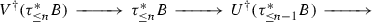in $${\text {DSec}}_n(I,\mathcal {C})$$, where $$U^\dagger$$ is the adjoint functor of Proposition 8.4, and it induces a long exact sequence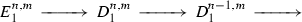This turns $$\langle D_1^{\cdot ,\cdot },E_1^{\cdot ,\cdot } \rangle$$ into an exact couple, and the corresponding spectral sequence converges to $${\text {Hom}}^\cdot (A,B)$$. To deduce (8.27), note that we have

\begin{aligned} E_1^{n,m} \cong {\text {Hom}}\left( V_\dagger \tau _{\le n}^*A,V^\dagger \tau _{\le n}^*B\right) \end{aligned}

by adjunction, and use the definition of the functors (8.26). $$\square$$

### Example 8.12

Take $$I= \cong ^o$$, with $$I=I_M$$ and the degree function as in Example 7.22. Then as in Example 4.13, a good stable model prefibration $$\langle \mathcal {C},\mathcal {C}' \rangle$$ over I is given by its fibers $$\langle \mathcal {C}_0,\mathcal {C}_0' \rangle$$, $$\langle \mathcal {C}_1,\mathcal {C}_1' \rangle$$ and a stable right-derivable functor $$\Phi :\langle \mathcal {C}_0,\mathcal {C}_0' \rangle \rightarrow \langle \mathcal {C}_0,\mathcal {C}_0' \rangle$$. Moreover, by Proposition 7.26 and Example 4.13, we have $${\text {DSec}}(I,\mathcal {C}) \cong {\text {Ho}}({\text {Sec}}(I,\mathcal {C})) \subset {\text {Ho}}({\text {Sec}}(I,\mathcal {C}')) \cong {\text {Ho}}(\mathsf{R}(\Phi ))$$, so that an object $$E \in {\text {DSec}}(I,\mathcal {C})$$ defines objects $$E_0 \in {\text {Ho}}(\mathcal {C}_0)$$, $$E_1 \in {\text {Ho}}(E_1)$$ and a morphism $$\alpha (E):E_1 \rightarrow R^\cdot \Phi E_0$$. Then by Lemma 3.16, we have $$\overline{L}_i(E) \cong E_i$$, $$i=0,1$$, $$\overline{M}_0(E) \cong E_0$$, and $$\overline{M}_1(E)$$ fits into a distinguished triangle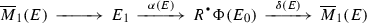(8.28)

The spectral sequence of Corollary 8.11 reduces to a complex with two terms, $${\text {Hom}}^\cdot (A_0,B_0)$$ and $${\text {Hom}}^\cdot (A_1,\overline{M}_1(B))$$, and the differential is the composition of the map $$R^\cdot \Phi :{\text {Hom}}^\cdot (A_0,B_0) \rightarrow {\text {Hom}}^\cdot (R^\cdot \Phi (A_0),R^\cdot \Phi (B_0))$$ and the map

\begin{aligned} {\text {Hom}}^\cdot (R^\cdot \Phi (A_0),R^\cdot \Phi (B_0)) \rightarrow {\text {Hom}}^{\cdot -1}(A_1,\overline{M}_1(B)) \end{aligned}

sending a map $$f:R^\cdot \Phi (A_0) \rightarrow R^\cdot \Phi (B_0)$$ to $$\delta (B) \circ f \circ \alpha (A)$$.

The spectral sequence of Corollary 8.11 is obviously functorial in A and B. Moreover, assume given another Reedy category $$I'$$ and a Reedy functor $$\gamma :I' \rightarrow I$$ that preserves the degrees. Then the spectral sequence is also functorial with respect to the pullback functor (8.3) in the following sense. For any $$n \ge 0$$, $$\gamma$$ induces a functor $$\gamma :I'_{\le n} \rightarrow I_{\le n}$$, we have $$\tau _{\le n} \circ \gamma ^* \cong \gamma ^* \circ \tau _{\le n}$$ and $$U \circ \gamma ^* \cong \gamma ^* \circ U$$, and then by adjunction, we also have natural maps $$V_\dagger \circ \gamma ^* \rightarrow \gamma ^* \circ V_\dagger$$, $$\gamma ^* \circ V^\dagger \rightarrow V^\dagger \circ \gamma ^*$$ that provide functorial maps

\begin{aligned} \overline{L}_i \circ \gamma ^* \rightarrow \overline{L}_{\gamma (i)}, \qquad \overline{M}_{\gamma (i)} \rightarrow \overline{M}_i \circ \gamma ^* \end{aligned}
(8.29)

after evaluation at an object $$i \in \overline{I'}_n$$. For any $$A,B \in {\text {DSec}}(I,\mathcal {C})$$, these maps provide a natural map

\begin{aligned} {\text {Hom}}(\overline{L}_{\gamma (i)}(A),\overline{M}_{\gamma (i)}(B)) \rightarrow {\text {Hom}}(\overline{L}_i(\gamma ^*A),\overline{M}_i(\gamma ^*B)), \end{aligned}

and collecting these maps together, we obtain a natural map between the $$E_1$$-terms of the spectral sequences for $${\text {Hom}}^\cdot (A,B)$$ and $${\text {Hom}}^\cdot (\gamma ^*A,\gamma ^*B)$$. In the $$E_\infty$$-term, this map corresponds to the pullback map $$\gamma ^*:{\text {Hom}}^\cdot (A,B) \rightarrow {\text {Hom}}^\cdot (\gamma ^*A,\gamma ^*B)$$.

To use effectively the spectral sequence of Corollary 8.11, we need some way to control the functors $$\overline{L}_i$$, $$\overline{M}_i$$, $$i \in I$$. It is convenient to introduce the following.

### Definition 8.13

For any object i in a Reedy category I, the completed latching category $$\overline{L}(i)$$ is the category of objects $$i' \in I$$ equipped with a map $$l:i' \rightarrow i$$ in the class L, and the completed matching category $$\overline{M}(i)$$ is the category of objects $$i' \in I$$ equipped with a map $$m:i \rightarrow i'$$ in the class M.

By definition, $$\overline{M}(i) \cong M(i)^<$$ is obtained by adding an initial object o to the usual matching category M(i), and $$o \in \overline{M}(i)$$ corresponds to the identity map $$\mathsf{id}:i \rightarrow i$$. Similarly, $$\overline{L}(i)^o \cong L(i)^{<o}$$, with the terminal object $$o \in \overline{L}(i)$$ corresponding to $$\mathsf{id}:i \rightarrow i$$. We also have natural forgetful functors

\begin{aligned} \lambda (i):\overline{L}(i) \rightarrow I, \qquad \mu (i):\overline{M}(i) \rightarrow I \end{aligned}
(8.30)

sending $$i' \rightarrow i$$ resp. $$i \rightarrow i'$$ to $$i' \in I$$. Moreover, the functors (8.17) and (8.22) extend to functors

\begin{aligned} \widetilde{\lambda }(i):{\text {DSec}}_{\le n}(I,\mathcal {C}) \rightarrow {\text {Ho}}(\overline{L}(i)), \quad \widetilde{\mu }(i):{\text {DSec}}_{\le n}(I,\mathcal {C}) \rightarrow {\text {Ho}}(\overline{M}(i)), \end{aligned}
(8.31)

with exactly the same definitions, and we still have the identification (8.23).

Now, for any finite ordered category J with an initial object $$o \in J$$, and any pointed model category $$\mathcal {C}$$, evaluation at o has a right-adjoint functor $$S:{\text {Ho}}(\mathcal {C}) \rightarrow {\text {Ho}}(\mathcal {C}^J)$$ sending $$E \in \mathcal {C}$$ to a functor $$E':J \rightarrow \mathcal {C}$$ such that $$E'(o)=E$$ and $$E'(j)=0$$ for $$j \ne o$$, and the functor S in turn has a right-adjoint functor $$S^\dagger :{\text {Ho}}(\mathcal {C}^J) \rightarrow {\text {Ho}}(\mathcal {C})$$. For a stable model pair $$\langle \mathcal {C},\mathcal {C}' \rangle$$, this induces a triangulated functor $$S^\dagger :{\text {Ho}}(\mathcal {C}^J) \rightarrow {\text {Ho}}(\mathcal {C})$$. Dually, we also have a functor $$T:{\text {Ho}}(\mathcal {C}) \rightarrow {\text {Ho}}(\mathcal {C}^{J^o})$$ left-adjoint to the evaluation at o, and it has a left-adjoint functor $$T_\dagger :{\text {Ho}}(\mathcal {C}^{J^o}) \rightarrow {\text {Ho}}(\mathcal {C})$$ and its triangulated version for stable model pairs. If $$J=$$, then these are exactly the functors (2.6), but they also exist for a more general category J. In particular, we can take $$J=\overline{M}(i)$$ or $$J=\overline{L}(i)^o$$, with the initial object o given by the identity arrow $$\mathsf{id}:i \rightarrow i$$ in both cases.

### Lemma 8.14

For any object i in a Reedy category I, any good stable model prefibration $$\langle \mathcal {C},\mathcal {C}' \rangle$$ over I, and any $$E \in {\text {DSec}}(I,\mathcal {C})$$, we have functorial identifications

\begin{aligned} \overline{L}_i(E) \cong T_\dagger (\widetilde{\lambda }(E)), \qquad \overline{M}_i(E) \cong S^\dagger (\widetilde{\mu }(E)). \end{aligned}

### Proof

Immediately follows from (8.18) resp. (8.25) applied both to I and to $$\overline{L}(i)$$ resp. $$\overline{M}(i)$$. $$\square$$

### Corollary 8.15

Assume given an object i in a Reedy category I and a good stable model prefibration $$\langle \mathcal {C},\mathcal {C}' \rangle$$ over I, and consider the natural maps

\begin{aligned} \overline{L}_o \circ \lambda (i)^* \rightarrow \overline{L}_i, \qquad \overline{M}_i \rightarrow \overline{M}_o \circ \mu (i)^* \end{aligned}
(8.32)

induced by (8.29), where $$\lambda (i)$$, $$\mu (i)$$ are as in (8.30), and o is the initial object $$\mathsf{id}:i \rightarrow i$$. Then both maps (8.32) are isomorphisms.

### Proof

We obviously have $$\widetilde{\lambda }(i) \cong \widetilde{\lambda }(o) \circ \lambda (i)$$ and $$\widetilde{\mu }(i) \cong \widetilde{\mu }(o) \circ \mu (i)$$. $$\square$$

For another useful corollary of Lemma 8.14, assume given a good stable model prefibration $$\langle \mathcal {C},\mathcal {C}' \rangle$$ over a Reedy category I, and a left-closed full subcategory $$I' \subset I$$ with the embedding functor $$\gamma :I' \rightarrow I$$, as in Lemma 8.9. Moreover, assume that the full embedding $$\gamma :I' \rightarrow I$$ admits a left-adjoint functor $$\gamma _\dagger :I \rightarrow I'$$, with the adjunction map $$a:\mathsf{Id}\rightarrow \gamma \circ \gamma _\dagger$$ compatible with the prefibration $$\mathcal {C}' \rightarrow I$$ in the sense of Definition 4.17.

### Corollary 8.16

In the assumptions above, the essential image of the fully faithful functor $$\gamma _*:{\text {DSec}}(I',\gamma ^*\mathcal {C}) \rightarrow {\text {DSec}}(I,\mathcal {C})$$ consists of derived sections that are homotopy cartesian along $$a(i):i \rightarrow \gamma (\gamma _\dagger (i))$$ for all $$i \in I$$.

### Proof

Since $$\gamma _*$$ is fully faithful, it suffices to prove that for any $$E \in {\text {DSec}}(I',\mathcal {C})$$, $$\gamma _*(E)$$ is homotopy cartesian along adjunction maps—then for any $$E' \in {\text {DSec}}(I,\mathcal {C})$$ homotopy cartesian along such maps, the adjunction map $$E' \rightarrow \gamma _*\gamma ^*E'$$ will be trivially an isomorphism. Definition 8.8 immediately implies that for any $$i \in I$$, the map a(i) is in M, and the functor $$\gamma _\dagger$$ sends maps in M to maps in M. Moreover, if we restrict $$\gamma _\dagger$$ to a functor $$\gamma _\dagger :\overline{M}(i) \rightarrow \overline{M}(\gamma _\dagger (i))$$, then it has a right-adjoint functor $$\gamma :\overline{M}(\gamma _\dagger (i)) \rightarrow \overline{M}(i)$$ sending $$m:\gamma _\dagger (i) \rightarrow i'$$ to the composition $$\gamma (m) \circ a$$. We need to prove that $$\gamma _*E$$ is homotopy cartesian along a(i), and by induction on $$\deg (i)$$, we may assume that for any non-trivial arrow $$i \rightarrow i'$$ representing an object in $$M(i) \subset \overline{M}(i)$$, E is homotopy cartesian along $$a(i')$$. Since a is compatible with the prefibration $$\mathcal {C}'$$, it is also compatible with the prefibration $${\text {Ho}}(\mathcal {C}) \rightarrow I$$, and then by (8.23), $$\widetilde{\mu }(i)(\gamma _*E)$$ is homotopy constant along maps $$a(i')$$. This means that the cone $$E'$$ of the adjunction map

\begin{aligned} \widetilde{\mu }(i)(\gamma _*E) \rightarrow \gamma _\dagger ^*\gamma ^*\widetilde{\mu }(i)(\gamma _*E) \end{aligned}
(8.33)

is supported at the initial object $$o \in M(i)$$, in the sense that $$E'(i') = 0$$ for any other object. We need to prove that $$E'(o) = 0$$ as well. If i lies in $$I' \subset I$$, $$a(i)=\mathsf{id}$$, so we may assume that this is not the case. Then $${\text {Ho}}(\mathcal {C}_i) \subset {\text {DSec}}(I,\mathcal {C})$$ lies in the kernel of the functor $$\gamma ^*$$, so that by adjunction and Lemma 8.14, we have $$S^\dagger (\widetilde{\mu }(i)(\gamma _*E)) = \overline{M}_i(\gamma _*E) = 0$$. On the other hand, since o does not lie in $$\gamma (\overline{M}(\gamma _\dagger (i))) \subset \overline{M}(i)$$, we have $$\gamma ^* \circ S = 0$$, so that $$S^\dagger \circ \gamma _\dagger ^* = 0$$ by adjunction, and the target of the map (8.33) also vanishes after we apply $$S^\dagger$$. Thus $$S^\dagger (E')=0$$, and since $$E'$$ is supported at o, this proves that $$E'=0$$. $$\square$$

### Functoriality

Another corollary of Proposition 8.4 is extended functoriality for the categories of derived sections. Namely, assume given a Reedy category I and two good stable model prefibrations $$\langle \mathcal {C}_0,\mathcal {C}_0' \rangle$$, $$\langle \mathcal {C}_1,\mathcal {C}_1' \rangle$$ over I.

### Definition 8.17

A stable morphism $$\Phi$$ from $$\langle \mathcal {C}_0,\mathcal {C}_0' \rangle$$ to $$\langle \mathcal {C}_1,\mathcal {C}_1' \rangle$$ is a functor $$\Phi :\mathcal {C}_0' \rightarrow \mathcal {C}_1'$$ over I such that for any object $$i \in I$$, $$\Phi (i)$$ is a stable right-derivable functor in the sense of Definition 3.2.

Note that we do not require that $$\Phi$$ is right-derivable globally over I in any sense. Nevertheless, it turns out that what we do require in Definition 8.17 is enough to construct a natural triangulated functor

\begin{aligned} R^\cdot \Phi :{\text {DSec}}(I,\mathcal {C}_0) \rightarrow {\text {DSec}}(I,\mathcal {C}_1). \end{aligned}
(8.34)

Namely, consider the prefibration $$\widetilde{\mathcal {C}}' \rightarrow I \times ^o$$ associated to $$\Phi$$ as in Example 4.14. By definition, the fibers of the projection $$\widetilde{\mathcal {C}}' \rightarrow ^o$$ over $$0,1 \in ^o$$ are the categories $$\mathcal {C}'_0$$ resp. $$\mathcal {C}'_1$$. Let $$\widetilde{\mathcal {C}} \subset \widetilde{\mathcal {C}}'$$ be the full subcategory spanned by $$\mathcal {C}_0 \subset \mathcal {C}'_0$$ and $$\mathcal {C}_1 \subset \mathcal {C}'_1$$. Equip $$I \times ^o$$ with the product of the Reedy structure on I and the standard Reedy structure on $$^o$$ such that all maps are in M, with the degree function $$\deg (i \times x) = \deg (i) + x$$, $$x = 0,1 \in ^o$$.

### Lemma 8.18

The pair $$\langle \widetilde{\mathcal {C}},\widetilde{\mathcal {C}}' \rangle$$ is a good stable model prefibration over the Reedy category $$I \times ^o$$.

### Proof

Since $$^o_L$$ is discrete, we have $$(I \times ^o)_L = (I_L \times 0) \sqcup (I_L \times 1)$$, so that Definition 7.12 (i) for $$\widetilde{\mathcal {C}}'$$ immediately follows from the corresponding properties of $$\mathcal {C}'_0$$ and $$\mathcal {C}'_1$$. For Definition 7.12 (ii), denote by g the only non-trivial map in $$^o$$. Then by definition, for any map $$f:i' \rightarrow i$$ in I, the transition functor $$(f \times g)^*$$ of the prefibration $$\widetilde{\mathcal {C}}'$$ is given by

\begin{aligned} (f \times g)^* \cong f^* \circ \Phi (i) \cong (f \times \mathsf{id})^* \circ (\mathsf{id}\times g)^*. \end{aligned}

This implies Definition 7.12 (ii), so that $$\widetilde{\mathcal {C}}' \rightarrow I \times ^o$$ is good. The rest immediately follows from Definition 8.17. $$\square$$

By Lemma 8.18, we have the triangulated category $${\text {DSec}}(I \times ^o,\widetilde{\mathcal {C}})$$. We also have Reedy functors $$s,t:I \rightarrow I \times ^o$$ given by $$s(i) = i \times 0$$, $$t(i) = i \times 1$$, both are left-closed embeddings in the sense of Definition 8.8, and we have $$s^*\widetilde{\mathcal {C}} = \mathcal {C}_0$$, $$t^*\widetilde{\mathcal {C}}= \mathcal {C}_1$$. By Lemma 8.9, the pullback functors $$s^*$$ and $$t^*$$ admit fully faithful right-adjoint functors $$t_*$$, $$s_*$$. Moreover, $$I \times 0 \subset I \times ^o$$ actually satisfies the stronger assumption of Lemma 8.10, so that we have a semiorthogonal decomposition

\begin{aligned} {\text {DSec}}(I \times ^o,\widetilde{\mathcal {C}}) = \langle t_*({\text {DSec}}(I,\mathcal {C}_1)),s_*({\text {DSec}}(I,\mathcal {C}_0)) \rangle . \end{aligned}
(8.35)

The functor (8.34) that we want to construct then emerges as the gluing functor of this semiorthogonal decomposition – namely, we can put

\begin{aligned} R^\cdot \Phi = t^* \circ s_*:{\text {DSec}}(I,\mathcal {C}_0) \rightarrow {\text {DSec}}(I,\mathcal {C}_1). \end{aligned}
(8.36)

If $$I = \mathsf{pt}$$ is a point category, then $$\widetilde{\mathcal {C}}' = \mathsf{R}(\Phi )$$, and $$t^* \circ s_*$$ coincides with the derived functor $$R^\cdot \Phi$$ by Lemma 3.16, so that the notation is consistent. For any Reedy functor $$\gamma :I' \rightarrow I$$, we have the natural adjunction map

\begin{aligned} \gamma ^* \circ R^\cdot \Phi \rightarrow R^\cdot \gamma ^*(\Phi ) \circ \gamma ^*. \end{aligned}
(8.37)

Moreover, the embedding s is right-adjoint to the projection $$I \times ^o \rightarrow I$$, and the adjunction map is compatible with the prefibration $$\widetilde{\mathcal {C}}$$. Thus the essential image of the functor $$s_*$$ is described in Corollary 8.16, and it is clear from the description that $$\gamma ^*$$ preserves this image. Therefore the map (8.37) is actually an isomorphism. In particular, for any object $$i \in I$$ and derived section $$E \in {\text {DSec}}(I,\mathcal {C})$$, we have $$\mathsf{ev}_i(R^\cdot \Phi (E)) \cong R^\cdot \Phi (\mathsf{ev}_i(E))$$.

Another useful compatibility is with the spectral sequences of Corollary 8.11. Namely, the functor (8.36) obviously preserves filtration by degree and sends $${\text {Ho}}(\mathcal {C}_{0,i}) \subset {\text {DSec}}(I,\mathcal {C}_0)$$, $$i \in I$$ into $${\text {Ho}}(\mathcal {C}_{1,i}) \subset {\text {DSec}}(I,\mathcal {C}_1)$$. Therefore by adjunction, we have natural maps

\begin{aligned} \overline{L}_i \circ R^\cdot \Phi \rightarrow R^\cdot \Phi (i) \circ \overline{L}_i, \qquad R^\cdot \Phi (i) \circ \overline{M}_i \rightarrow \overline{M}_i \circ R^\cdot \Phi , \end{aligned}
(8.38)

and by (8.18) and (8.25), these maps are isomorphisms. Then for any $$A,B \in {\text {DSec}}(I,\mathcal {C}_0)$$, $$i \in I$$, the functor $$R^\cdot \Phi (i)$$ induces a map

\begin{aligned} {\text {Hom}}^\cdot (\overline{L}_i(A),\overline{M}_i(B)) \rightarrow {\text {Hom}}(\overline{L}_i(R^\cdot \Phi (A)),\overline{M}_i(R^\cdot \Phi (B)), \end{aligned}

and together, these maps define a map between the $$E_1$$-terms of the spectral sequences of Corollary 8.11. In the $$E_\infty$$-term, this corresponds to the map given by the functor $$R^\cdot \Phi$$.

We can also combine this construction with Example 8.12 to apply it to derived sections over the category $$I \times ^o$$. Namely, any good stable model prefibration $$\langle \widetilde{\mathcal {C}},\widetilde{\mathcal {C}}' \rangle$$ over $$I \times ^o$$ such that $$\widetilde{\mathcal {C}}' \rightarrow ^o$$ is also a prefibration defines by restriction good stable model prefibrations $$\langle \mathcal {C}_0,\mathcal {C}_0' \rangle$$, $$\langle \mathcal {C}_1,\mathcal {C}_1' \rangle$$ over I, and a stable morphism $$\Phi :\langle \mathcal {C}_0,\mathcal {C}_0' \rangle \rightarrow \langle \mathcal {C}_1,\mathcal {C}_1' \rangle$$, while every derived section $$E \in {\text {DSec}}(I \times ^o,\widetilde{\mathcal {C}})$$ gives derived sections $$E_0 \in {\text {DSec}}(I,\mathcal {C}_0)$$, $$E_1 \in {\text {DSec}}(I,\mathcal {C}_1)$$ and a morphism $$\alpha (E):E_1 \rightarrow R^\cdot \Phi E_0$$. By Corollary 8.15, we have

\begin{aligned} \overline{L}_{i \times 0} \cong \overline{L}_i \circ s^*, \quad \overline{M}_{i \times 0} \cong \overline{M}_i \circ s^*, \quad \overline{L}_{i \times 1} \cong t^* \circ \overline{L}_i \end{aligned}
(8.39)

for any object $$i \in I$$. Moreover, the adjoint functor $$t_*$$ of (8.35) itself admits a right-adjoint functor $$t^!$$ provided by the semiorthogonal decomposition (8.35), and for any $$E \in {\text {DSec}}(I \times ^o,\widetilde{\mathcal {C}})$$, we have a distinguished triangle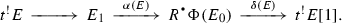(8.40)

Then for any $$i \in I$$, $${\text {Ho}}(\widetilde{C}_{i \times 1}) \subset {\text {DSec}}(I \times ^o,\widetilde{\mathcal {C}})$$ lies in the essential image of the fully faithful functor $$t_*$$, so that by adjunction, we have an isomorphism

\begin{aligned} \overline{M}_{i \times 1} \cong \overline{M}_i \circ t^!. \end{aligned}
(8.41)

Now for any $$A,B \in {\text {DSec}}(I \times ^o,\widetilde{\mathcal {C}})$$, we can combine (8.39) and (8.41) and obtain a natural map

\begin{aligned} {\text {Hom}}^\cdot (R^\cdot \Phi (i)\overline{L}_{i \times 0}(A),R^\cdot \Phi (i)\overline{M}_{i \times 0}(B)) \rightarrow {\text {Hom}}^{\cdot -1}(\overline{L}_{i \times 1}(A),\overline{M}_{i \times 1}(B)) \end{aligned}

sending a map f to $$\delta (B) \circ f \circ \alpha (A)$$, as in Example 8.12. Composing this map with the map induced by the functor $$R^\cdot \Phi (i)$$, and taking the sum over all objects $$i \in I$$, we obtain a map

\begin{aligned} E_1(A_0,B_0) \rightarrow E_1(A_1,\overline{B}_1), \end{aligned}
(8.42)

where $$E_1(-,-)$$ stands for the $$E_1$$-term of the spectral sequence of Corollary 8.11, and $$\overline{B}_1 = t^!(B)$$.

### Lemma 8.19

The map (8.42) is compatible with the differentials, and the $$E_1$$-term $$E_1(A,B)$$ of the spectral sequence of Corollary 8.11 with its differential is the cone of this map.

### Proof

The distinguished triangle (8.40) for the object B is induced by a distinguished triangle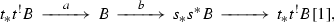(8.43)

where a and b are the adjunction maps. Since $$E_1(A,B)$$ is functorial in B, this induces a short exact sequence of complexes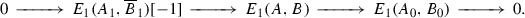Moreover, if we forget the differentials, that this sequence is canonically split, so that $$E_1(A,B)$$ is the cone of some map from $$E_1(A_0,B_0)$$ to $$E_1(A_1,\overline{B}_1)$$. Checking that the map indeed coincides with (8.42) can be done separately for each of the terms $${\text {Hom}}(\overline{L}_i(A_0),\overline{M}_i(B_0))$$, $$i \in I$$ of (8.27). Then by functoriality and induction on degree, we may assume that $$I = I_{\le n}$$, $$n = \deg (i)$$, and moreover, that B vanishes after restriction to $$I_{\le n-1} \times ^o$$. But in this case, since the embedding $$I_{\le n-1} \times ^o \subset I_{\le n} \times ^o$$ obviously satisfies the assumptions of Lemma 8.10, the claim reduces to the case $$I=\mathsf{pt}$$ considered in Example 8.12. $$\square$$

## General gluing

We can now combine the homotopical results of Sects. 7 and 8, on one hand, and the simplicial technology of Sects. 5 and 6, on the other hand. The result is a derived version of the gluing constructions of Sect. 4.5. In this section, we treat the case of cosections, with comonads and coalgebras postponed until Sect. 10. We define the notion of a stable model family over a category I, our version of a family of enhanced triangulated categories, and for any such family $$\mathcal {C}$$, we construct the triangulated category $${\text {DRec}}(I,\mathcal {C})$$ of derived cosections. We show that $${\text {DRec}}(I,\mathcal {C})$$ has the same basic functoriality as the usual category of cosections of Definition 4.21. We then construct a spectral sequence that refines the isomorphism of Lemma 5.13 and gives one some control over $${\text {DRec}}(I,\mathcal {C})$$. As an application, we show that a triangulated category coming from a stable model pair is automatically enriched over the stable homotopy category, and prove additional results on functoriality in the case of a constant family $$\mathcal {C}= \mathcal {C}_0 \times I$$, when $${\text {DRec}}(I,\mathcal {C})$$ is the enhanced version of the category of functors from I to $${\text {Ho}}(\mathcal {C}_0)$$. In particular, we construct the left and right-derived Kan extension functors.

### Stable model families

Let us apply the technology of Reedy model structures to special prefibrations over categories of simplices in the sense of Definition 5.1.

Recall that for any simplicial set X, the category of simplices $$\Delta X$$ carries a natural Reedy structure of Example 7.8. The opposite category $$(\Delta X)^o$$ is then also a Reedy category, with the opposite Reedy structure.

### Lemma 9.1

A prefibration $$\mathcal {C}\rightarrow (\Delta X)^o$$ special in the sense of Definition 5.10 is good in the sense of Definition 7.12 if and only if it is normalized.

### Proof

Every surjective map in $$\Delta$$ is special, so that Definition 7.12 (i) follows from Definition 5.10, and Definition 7.12 (ii) is then precisely equivalent to the normalization condition. $$\square$$

### Definition 9.2

For any simplicial set X, a good stable model prefibration $$\langle \mathcal {C},\mathcal {C}' \rangle$$ over the category $$(\Delta X)^o$$ is homotopy special if the corresponding prefibration (8.1) is special in the sense of Definition 5.10. A derived section $$E \in {\text {DSec}}((\Delta X)^o,\mathcal {C})$$ of such a stable model prefibration is homotopy special if the section $$\mathsf{ev}(E)$$ obtained by applying the evaluation functor (8.5) is special in the sense of Definition 5.10.

For any homotopy special good stable model prefibration $$\langle \mathcal {C},\mathcal {C}' \rangle$$ over $$(\Delta X)^o$$, we denote by $${\text {DSec}}_+((\Delta X)^o,\mathcal {C}) \subset {\text {DSec}}((\Delta X)^o,\mathcal {C})$$ the full subcategory spanned by homotopy special sections. It is obviously triangulated, and comes from a natural stable model pair. The evaluation functor (8.5) induces a functor

\begin{aligned} \mathsf{ev}:{\text {DSec}}_+((\Delta X)^o,\mathcal {C}) \rightarrow {\text {Sec}}_+((\Delta X)^o,{\text {Ho}}(\mathcal {C}/(\Delta X)^o)). \end{aligned}
(9.1)

The functor is of course not an equivalence, and the category on the right-hand side is not triangulated—it is only the category of sections of a prefibration with triangulated fibers. The only remnant of the triangulated structure that survives in such a category is homological shift: the transition functors of the prefibration $${\text {Ho}}(\mathcal {C}/(\Delta X)^o)$$ commute with shifts, so that we have a global endofunctor $$X \mapsto X$$ on $${\text {Sec}}_+((\Delta X)^o,{\text {Ho}}(\mathcal {C}/(\Delta X)^o))$$. The evaluation functor (9.1) commutes with this functor.

An abundant source of normalized special prefibrations over categories of simplices is provided by Proposition 6.11. To incorporate model structures into the picture, we introduce the following.

### Definition 9.3

A model family $$\mathcal {C}$$ over a small category I is a category $$\mathcal {C}$$ equipped with a precofibration $$\mathcal {C}\rightarrow I$$ and classes of fiberwise maps C, F, W such that for every $$i \in I$$, the fiber $$\mathcal {C}_i$$ with the classes C, F, W is a model category, and for every map $$f:i \rightarrow i'$$ in I, the transition functor $$f_!:\mathcal {C}_i \rightarrow \mathcal {C}_{i'}$$ is right-derivable. A stable model family over I is a model family $$\mathcal {C}' \rightarrow I$$ equipped with a full subcategory $$\mathcal {C}\subset \mathcal {C}'$$ such that for any $$i \in I$$, $$\langle \mathcal {C}_i,\mathcal {C}'_i \rangle$$ is a stable model pair, and for any map $$f:i \rightarrow i'$$, the functor $$f_!$$ is stable.

Then for any model family $$\mathcal {C}\rightarrow I$$, the barycentric expansion $$S(I,\mathcal {C}) \rightarrow \Delta I$$ of Definition 6.9 is a model prefibration in the sense of Definition 7.15. Moreover, by Lemma 9.1, its reflection $$S(I,\mathcal {C})^\flat$$ of Definition 5.12 is good in the sense of Definition 7.12. Recall that explicitly, the fiber $$S(I,\mathcal {C})^\flat _{\langle [n],\kappa \rangle }$$ over an object $$\langle [n],\kappa \rangle \in (\Delta I)^o$$ is naturally identified with the fiber $$\mathcal {C}_{\kappa (n)}$$. For a stable model family $$\langle \mathcal {C},\mathcal {C}' \rangle$$, we have a good model prefibration $$\widetilde{\mathcal {C}'} = S(I,\mathcal {C}')^\flat \rightarrow (\Delta I)^o$$, and if we let $$\widetilde{\mathcal {C}} \subset \widetilde{\mathcal {C}}'$$ be the full subcategory spanned by the subcategories $$\mathcal {C}_{\kappa (n)} \subset \mathcal {C}'_{\kappa (n)} \cong \widetilde{\mathcal {C}}'_{\langle [n],\kappa \rangle }$$, then $$\langle \widetilde{\mathcal {C}},\widetilde{\mathcal {C}'} \rangle$$ is obviously a good stable model prefibration over $$\Delta I$$ homotopy special in the sense of Definition 9.2.

### Definition 9.4

Assume given a stable model family $$\langle \mathcal {C},\mathcal {C}' \rangle$$ over a small category I. Then the category $${\text {DRec}}(I,\mathcal {C})$$ of derived cosections of the family $$\langle \mathcal {C},\mathcal {C}' \rangle$$ is given by

\begin{aligned} {\text {DRec}}(I,\mathcal {C}) = {\text {DSec}}_+((\Delta I)^o,\widetilde{\mathcal {C}}), \end{aligned}

where $$\langle \widetilde{\mathcal {C}},\widetilde{\mathcal {C}}' \rangle$$ is the corresponding homotopy special good stable model prefibration over $$(\Delta I)^o$$.

The category of derived cosections is obviously functorial in I—for any functor $$\gamma :I' \rightarrow I$$ from another small category $$I'$$, $$\langle \gamma ^*,\gamma ^*\mathcal {C}' \rangle$$ is a stable model family, and we have the triangulated pullback functor

\begin{aligned} \gamma ^*:{\text {DRec}}(I,\mathcal {C}) \rightarrow {\text {DRec}}(I',\gamma ^*\mathcal {C}). \end{aligned}
(9.2)

For any stable model family $$\langle \mathcal {C},\mathcal {C}'\rangle$$ over I, the categories $${\text {Ho}}(\mathcal {C}_i)$$, $$i \in I$$ with the transition functors $$R^\cdot f_!$$ form a precofibration over I that we will denote by $${\text {Ho}}(\mathcal {C}/I)$$. Then we have $$S(I,{\text {Ho}}(\mathcal {C}/I))^\flat \cong {\text {Ho}}(\widetilde{\mathcal {C}})$$, and the evaluation functor (9.1) induces a natural functor

\begin{aligned} \mathsf{ev}:{\text {DRec}}(I,\mathcal {C}) \rightarrow {\text {Rec}}(I,{\text {Ho}}(\mathcal {C}/I)). \end{aligned}
(9.3)

It is our contention that it is $${\text {DRec}}(I,\mathcal {C})$$ that should be thought of as the correct derived gluing of the family $$\mathcal {C}$$. To illustrate this contention, let us show that the categories of derived cosections admits a version of the equivalence (4.31).

Assume given a small category I and a stable model family $$\langle \mathcal {C},\mathcal {C}' \rangle$$ over the category $$I^<$$. As in (4.31), we have the restriction $$\langle j^*\mathcal {C},j^*\mathcal {C}' \rangle$$ of the family $$\langle \mathcal {C},\mathcal {C}' \rangle$$ to $$I \subset I^<$$, a fiber $$\langle \mathcal {C}_o,\mathcal {C}'_o \rangle$$ over $$o \in I^<$$, and a functor $$\Phi :\mathcal {C}'_o \rightarrow {\text {Rec}}(I,j^*\mathcal {C}') \cong {\text {Sec}}_+((\Delta I)^o,S(I,\mathcal {C}')^\flat )$$. The functor $$\Phi$$ defines a stable morphism

\begin{aligned} \Phi :\mathcal {C}'_o \times (\Delta I)^o \rightarrow S(I,\mathcal {C}')^\flat \end{aligned}

in the sense of Definition 8.17, and this stable morphism is homotopy cartesian over special maps. Then (8.36) together with the pullback functor $$\tau ^*$$ with respect to the tautological projection $$\tau :(\Delta I^<)^o \rightarrow \mathsf{pt}$$ provide a triangulated functor

\begin{aligned} R^\cdot \Phi \circ \tau ^*:{\text {Ho}}(\mathcal {C}_0) \rightarrow {\text {DRec}}(I,I \times \mathcal {C}_0) \rightarrow {\text {DRec}}(I,j^*\mathcal {C}). \end{aligned}
(9.4)

### Lemma 9.5

In the assumptions above, the pullback functor $$o^*$$ resp. $$j^*$$ of (9.2) admits a fully faithful left resp. right-adjoint functor, and we have a semiorthogonal decomposition

\begin{aligned} {\text {DRec}}(I^<,\mathcal {C}) = \langle {\text {Ho}}(\mathcal {C}_o),{\text {DRec}}(I,j^*\mathcal {C}) \rangle \end{aligned}
(9.5)

in the sense of Definition 3.15 whose gluing functor is the functor (9.4).

### Proof

The embeddings $$\{o\} \rightarrow I^<$$, $$j:I \rightarrow I^<$$ define fully faithful embeddings of simplicial replacements $$i_0:\Delta \rightarrow \Delta I^<$$, $$i_1:\Delta I \rightarrow \Delta I^<$$. Denote by $$(\Delta I^<)_0,(\Delta I^<)_1 \subset \Delta I^<$$ the complements to the images of these embeddings (that is, the full subcategories spanned by objects not in the image), and say a map in any of these categories is special if it is special in $$\Delta I^<$$. Then the embedding $$i_0^o:\Delta ^o \rightarrow (\Delta I^<)^o$$ is left-closed in the sense of Definition 8.8 and satisfies the assumptions of Lemma 8.10. Therefore we have a semiorthogonal decomposition

\begin{aligned} {\text {DSec}}((\Delta I^<)^o,\widetilde{\mathcal {C}}) = \langle {\text {DSec}}(\Delta ^o,\widetilde{\mathcal {C}}),{\text {DSec}}((\Delta I^<)_0^o,\widetilde{\mathcal {C}})\rangle , \end{aligned}
(9.6)

where $$\widetilde{\mathcal {C}}$$ on the left is as in Definition 9.4, and by abuse of notation, $$\widetilde{\mathcal {C}}$$ on the right stands for its restrictions to $$\Delta ^o,(\Delta I^<)_0^o \subset (\Delta I^<)$$. Say that a derived section of $$\widetilde{\mathcal {C}}$$ on either of these two categories is homotopy special if it is homotopy cartesian along all special maps, and let $${\text {DSec}}_+ \subset {\text {DSec}}$$ stand for the full subcategory spanned by homotopy special sections. Then the restriction functors send homotopy special sections to homotopy special sections, so that (9.6) induces a semiorthogonal decomposition

\begin{aligned} {\text {DRec}}(I^<,\mathcal {C}) = \langle {\text {DSec}}_+(\Delta ^o,\widetilde{\mathcal {C}}),{\text {DSec}}_+((\Delta I^<)_0^o,\widetilde{\mathcal {C}})\rangle . \end{aligned}
(9.7)

The embedding $$\gamma :\mathsf{pt}\rightarrow \Delta ^o$$ onto $$ \in \Delta ^o$$ is a left-closed embedding satisfying the assumptions of Corollary 8.16, so that the image of the full embedding $$\gamma _*:{\text {Ho}}(\mathcal {C}_o) \rightarrow {\text {DSec}}(\Delta ^o,\widetilde{\mathcal {C}})$$ is described in Corollary8.16, and one immediately checks that it consists of homotopy special sections, so that $${\text {DSec}}_+(\Delta ^o,\widetilde{\mathcal {C}})$$ is equivalent to $${\text {Ho}}(\mathcal {C}_o)$$. On the other hand, the embedding $$i_1:\Delta I \rightarrow \Delta I^<$$ factors through an embedding $$\overline{i}_1:\Delta I \rightarrow (\Delta I)^<_0$$, and $$\overline{i}^o_1$$ is also a left-closed embedding satisfying the assumptions of Corollary 8.16; in this case, it shows that the restriction functor

\begin{aligned} \overline{i}_1^*:{\text {DSec}}_+((\Delta I^<)^o_0,\widetilde{\mathcal {C}}) \rightarrow {\text {DSec}}_+((\Delta I)^o,\widetilde{\mathcal {C}}) \cong {\text {DRec}}(I,j^*\mathcal {C}) \end{aligned}

is an equivalence. With these identifications, (9.7) is exactly (9.5). To compute the gluing functor, note that $$i_0:\Delta \rightarrow \Delta I^<$$ also factors through an embedding $$\overline{i}_0:\Delta \rightarrow (\Delta I^<)_1$$, and apply Corollary 8.16 to the opposite embedding $$\overline{i}_0^o$$. $$\square$$

### The standard differential

Let us now show that for homotopy special sections A, B of homotopy special good stable model prefibrations, one can actually compute the first differential and the $$E_2$$-term of the spectral sequence of Corollary 8.11. Recall that a simplex $$x \in X([n])$$ in a simplicial set X is non-degenerate if it does not lie in the image of the map $$X(f):X([n']) \rightarrow X([n])$$ for a non-identical surjective map $$f:[n] \rightarrow [n']$$.

### Lemma 9.6

Assume given a simplicial set X and a homotopy special good stable model prefibration $$\langle \mathcal {C},\mathcal {C}' \rangle$$ over the category $$(\Delta X)^o$$. Then for any $$E \in {\text {DSec}}_+((\Delta X)^o,\mathcal {C})$$ and $$\langle [n],x \rangle \in \Delta X$$, we have

\begin{aligned} \overline{L}_{\langle [n],x \rangle }(E) \cong \mathsf{ev}_{\langle [n],x \rangle }(E) \end{aligned}
(9.8)

if the simplex $$x \in X([n])$$ is non-degenerate, and 0 otherwise.

### Proof

For any simplex $$x \in X([n])$$, there exists a unique non-degenerate simplex $$x' \in X([n'])$$ and surjective map $$f:[n] \rightarrow [n']$$ such that $$x = f^*x'$$. The completed latching category $$\overline{L}(\langle [n],x \rangle )$$ of Definition 8.13 is then naturally equivalent to the product $$^{n-n'}$$ of $$n-n'$$ copies of the category . If x itself is non-degenerate, this is just the point, and then Lemma 8.14 immediately provides the isomorphism (9.8). If not, then by Lemma 8.14, $$\overline{L}_{\langle [n],x \rangle }$$ can still be computed after restriction to $$\overline{L}(\langle [n],x \rangle ) \cong ^{n-n'}$$, and the corresponding functor $$\overline{L}_o$$ on the completed latching category is obtained by applying the functor $$T_\dagger$$ of (2.6) along each of the $$n-n'$$ coordinates. Since E is homotopy special, $$\lambda (\langle [n],x \rangle )^*E$$ sends any map in $$\overline{L}(\langle [n],x \rangle )$$ to a weak equivalence, and we obtain 0 already after the first application of $$T_\dagger$$. $$\square$$

Now assume given a simplicial set X, a homotopy special good stable model prefibration $$\langle \mathcal {C},\mathcal {C}' \rangle$$ over the category $$(\Delta X)^o$$, and two homotopy special derived sections $$A,B \in {\text {DSec}}_+((\Delta X)^o,\mathcal {C})$$. For any integer l, denote

\begin{aligned} {\mathcal {H}{} { om}}^l(A,B) = {\mathcal {H}{} { om}}(\mathsf{ev}(A),\mathsf{ev}(B[l])_\flat ):\Delta X \rightarrow {\text {Sets}}, \end{aligned}
(9.9)

where B[l] stands for the cohomological shift, $$\mathsf{ev}$$ is the functor (9.1), and $${\mathcal {H}{} { om}}(\mathsf{ev}(A),\mathsf{ev}(B)_\flat )$$ is as in Lemma 5.13. Explicitly, for any $$\langle [n],x \rangle \in \Delta X$$, we have

\begin{aligned} {\mathcal {H}{} { om}}^l(A,B)(\langle [n],x \rangle ) \cong {\text {Hom}}(\mathsf{ev}_{\langle [n],x \rangle }(A),R^\cdot s^{o*}\mathsf{ev}_{\langle ,s^*x \rangle }(B[l])), \end{aligned}
(9.10)

where $$s:\langle ,s^*x \rangle \rightarrow \langle [n],x \rangle$$ is the base special map of Remark 5.2. Since the categories $${\text {Ho}}(\mathcal {C}_{\langle [n],x \rangle })$$ are triangulated, the functors (9.9) actually take values in abelian groups. Recall that for any small category I and functor E from I to abelian groups, the cohomology groups $$H^\cdot (I,E)$$ are given by

\begin{aligned} H^\cdot (I,E) = R^\cdot \mathsf{lim}_I(E), \end{aligned}

where $$R^\cdot \mathsf{lim}_E$$ are the derived functors of the limit functor $$\mathsf{lim}_I$$.

### Proposition 9.7

In the assumptions above, the $$E_2$$-term $$E_2(A,B)$$ of the spectral sequence of Corollary 8.11 is given by

\begin{aligned} E_2(A,B) \cong H^\cdot (\Delta X,{\mathcal {H}{} { om}}^\cdot (A,B)), \end{aligned}
(9.11)

and the isomorphism is functorial in A, B and X.

### Proof

Recall that since $$\pi :\Delta X \rightarrow \Delta$$ is a discrete Grothendieck fibration, the cohomology $$H^\cdot (\Delta X,E)$$ with any coefficients can be computed by first applying the right Kan extension functor $$\pi _*$$ and then take the standard cochain complex of the cosimplicial abelian group $$\pi _*E$$. Explicitly, this gives the complex $$C_\cdot (E)$$ with terms

\begin{aligned} C^n(E) = \coprod _{x \in X([n])}E(\langle [n],x \rangle ) \end{aligned}
(9.12)

and the usual differential given by the alternating sum of the face maps. Moreover, it suffices to take the normalized cochain complex, so that the product in (9.12) is taken only over non-degenerate simplices $$x \in X([n])$$. By Lemma 9.6, the $$E_1$$-term $$E_1(A,B)$$ of the spectral sequence of Corollary 8.11 is also given by a product (8.27) of terms indexed by non-degenerate simplices. Comparing (9.10) and (8.27), we see that it suffices to construct isomorphisms

\begin{aligned} R^\cdot s^{o*}\mathsf{ev}_{\langle , s^*x \rangle }(B) \cong \overline{M}_{\langle [n],x \rangle }(B)[n] \end{aligned}
(9.13)

for all $$[n] \in \Delta$$ and non-degenerate $$x \in X([n])$$ that are functorial in B and X, and such that the induced isomorphism

\begin{aligned} C^\cdot ({\mathcal {H}{} { om}}^\cdot (A,B)) \cong E_1^{\cdot ,\cdot }(A,B) \end{aligned}
(9.14)

is compatible with the differentials.

By Lemma 8.14, to compute the matching functor $$\overline{M}_{\langle [n],x \rangle }$$ in (9.13), we can restrict the good stable model prefibration $$\langle \mathcal {C},\mathcal {C}' \rangle$$ to the completed matching category $$\overline{M}(\langle [n],x \rangle )$$. This category does not depend on x, nor on X, and is naturally opposite to the partially ordered set $$\overline{V}_n$$ of non-empty subsets $$S \subset [n]$$ in the ordinal [n]. It is convenient to add the empty subset o, consider the partially ordered set $$V_n \cong ^{n+1}$$ of all subsets in [n], and extend $$\langle \mathcal {C},\mathcal {C}' \rangle$$ to $$V_n^o$$ by setting $$\mathcal {C}_o=\mathcal {C}'_o=0$$. Restriction with respect to the embedding $$\overline{V}_n \subset V_n$$ induces a functor

\begin{aligned} {\text {DSec}}\left( \overline{V}_n^o,\mathcal {C}\right) \rightarrow {\text {DSec}}\left( V_n^o,\mathcal {C}\right) , \end{aligned}

and this functor is an equivalence by the same argument as in the proof of Lemma 8.6. But now we can consider the embedding $$s_n:[n-1] \rightarrow [n]$$ onto the initial segment of the ordinal [n], and for any $$S \subset [n]$$, the intersection $$S \cap [n-1]$$ is a subset in $$[n-1]$$. This gives an identification $$V_n \cong V_{n-1} \times $$, with $$V_{n-1} \times 0 \subset V_n$$ embedded via the embedding $$s_n$$, and puts us into the situation of Lemma 8.18. The distinguished triangle (8.40) then provides a distinguished triangle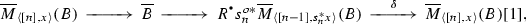where $$\overline{B}$$ is obtained by restricting B to $$V_{n-1}^0 \times 1 \subset V_n^o$$ and then applying the functor $$\overline{M}_{[n-1] \times 1}$$. Since B is homotopy special, this restriction is homotopy cartesian along all maps, so that $$\overline{B} = 0$$ as soon as $$n \ge 2$$ by the same argument as in Lemma 9.6. Thus the map $$\delta$$ in the triangle is an isomorphism, and by induction on n, we obtain the desired isomorphism (9.13).

The construction is obviously functorial in X and B, so to finish the proof, it remains to check that (9.14) is compatible with the differentials. However, since the spectral sequence of Corollary 8.11 is functorial with respect to base change, this can also be checked after restricting to $$\overline{M}(\langle [n],x \rangle )$$. Then it immediately follows from Lemma 8.19 by induction on n. $$\square$$

As an application of Proposition 9.7, let us show that for any stable model pair $$\langle \mathcal {C},\mathcal {C}' \rangle$$, the category $${\text {Ho}}(\mathcal {C})$$ is automatically enriched over the stable homotopy category, although in a rather weak sense. Fix a stable model pair $$\langle \mathcal {C},\mathcal {C}' \rangle$$. For any simplicial set X, consider the constant Grothendieck fibration $$\langle \mathcal {C}\times (\Delta X)^o,\mathcal {C}' \times (\Delta X)^o \rangle$$ over $$(\Delta X)^o$$, and denote

\begin{aligned} {\mathcal {D}}(X,\mathcal {C}) = {\text {DSec}}_+((\Delta X)^o,\mathcal {C}\times (\Delta X)^o). \end{aligned}

For any simplicial subset $$Y \subset X$$, denote by $${\mathcal {D}}(X,Y,\mathcal {C}) \subset {\mathcal {D}}(X,\mathcal {C})$$ the kernel of the restriction functor $${\mathcal {D}}(X,\mathcal {C}) \rightarrow {\mathcal {D}}(Y,\mathcal {C})$$. Since $$(\Delta Y)^o \subset (\Delta X)^o$$ is obviously a left-closed Reedy subcategory in the sense of Definition 8.8, we have a semiorthogonal decomposition $${\mathcal {D}}(X,\mathcal {C}) = \langle {\mathcal {D}}(X,Y,\mathcal {C}),{\mathcal {D}}(Y,\mathcal {C}) \rangle$$ provided by Lemma 8.9. If we also have a simplicial subset $$Z \subset Y$$, then this restricts to a semiorthogonal decomposition

\begin{aligned} {\mathcal {D}}(X,Z,\mathcal {C}) = \langle {\mathcal {D}}(X,Y,\mathcal {C}),{\mathcal {D}}(Y,Z,\mathcal {C}) \rangle . \end{aligned}
(9.15)

Any object $$E \in {\text {Ho}}(\mathcal {C})$$ defines a constant derived section $$E_X \in {\mathcal {D}}(X,\mathcal {C})$$. Projecting $$E_X$$ with respect to the semiorthogonal decomposition, we obtain a natural object $$E_{X,Y} \in {\mathcal {D}}(X,Y,\mathcal {C})$$, and for any triple $$Z \subset Y \subset X$$, we then have a natural distinguished triangle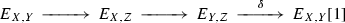(9.16)

in the triangulated category $${\mathcal {D}}(X,Z,\mathcal {C})$$. Now for any pair of simplicial sets $$Y \subset X$$ and two objects $$A,B \in {\text {Ho}}(\mathcal {C})$$, let

\begin{aligned} H^n(A,B)(X,Y) = {\text {Hom}}(A_X,B_{X,Y}[n]). \end{aligned}
(9.17)

For any triple $$Z \subset Y \subset X$$, we have $${\text {Hom}}(A_X,B_{Y,Z}) \cong {\text {Hom}}(A_Y,B_{Y,Z})$$ by adjunction, so that the connecting differential $$\delta$$ in (9.16) induces a functorial map

\begin{aligned} H^\cdot (A,B)(Y,Z) \rightarrow H^{\cdot +1}(A,B)(X,Y). \end{aligned}
(9.18)

### Lemma 9.8

For any $$A,B \in {\text {Ho}}(\mathcal {C})$$, the functors (9.17) together with the maps (9.18) form a generalized cohomology theory.

### Proof

All the axioms except for excision and homotopy invariance are obvious. For excision and homotopy invariance, use the spectral sequence of Corollary 8.11 to compute $$H^\cdot (A,B)(X,Y)$$, and note that the functor $${\mathcal {H}{} { om}}(A_X,B_X)$$ is actually constant for any simplicial set X, so that the right-hand side of (9.11) reduces to the ordinary relative cohomology $$H^\cdot (X,Y;-)$$. Thus by Proposition 9.7, both properties hold already in the $$E_2$$-term. $$\square$$

### Remark 9.9

By Lemma 9.8, for any $$A,B \in {\text {Ho}}(\mathcal {C})$$, we have a an object $$H^\cdot (A,B)$$ in the stable homotopy category, well-defined up to an isomorphism.

Of course, this is much weaker than even the usual categorical notion of enrichment—proving that would require defining $$H^\cdot (A,B)$$ up to a unique isomorphism and checking that the correspondence $$A,B \mapsto H^\cdot (A,B)$$ is compatible with compositions, and it does not seem possible to do either in terms of generalized cohomology theories.

### Specialization

Now assume given a model family $$\langle \mathcal {C},\mathcal {C}' \rangle$$ over a small category I, and assume that it satisfies two additional properties:

1. (i)

the projection $$\mathcal {C}' \rightarrow I$$ is a Grothendieck cofibration, and

2. (ii)

for any map $$f:i \rightarrow i'$$ in I, the transition functor $$f_!:\mathcal {C}'_i \rightarrow \mathcal {C}'_{i'}$$ preserves finite limits and sends $$\mathcal {C}_i \subset \mathcal {C}'_i$$ into $$\mathcal {C}_{i'} \subset \mathcal {C}'_{i'}$$.

Then by (i) and (ii), the induced functor $$\mathcal {C}\rightarrow I$$ is also a Grothendieck cofibration, and we then have $${\text {Rec}}(I,\mathcal {C}) \cong {\text {Sec}}(I^o,\mathcal {C}^\perp )$$. We also have $$S(I,\mathcal {C}) \cong \xi ^*\mathcal {C}^\flat$$, where $$\xi$$ is the functor (5.4), and $$S(I,\mathcal {C})^\flat \cong \beta ^{o*}\mathcal {C}^\perp$$, where $$\beta$$ is the functor (5.7). Moreover, we have $${\text {DSec}}((\Delta I)^o,\beta ^{o*}\mathcal {C}^\perp ) \cong {\text {Ho}}({\text {Sec}}((\Delta I)^o,\beta ^{o*}\mathcal {C}^\perp ))$$ by (ii) and Proposition 7.26, so that we do not need to consider matching expansions to describe derived sections of $$\beta ^{o*}\mathcal {C}^\perp$$.

As it turns out, if restrict our attention to homotopy special derived sections, then we do not even need to consider the simplicial expansion $$\Delta I$$.

### Proposition 9.10

Assume given a model family $$\langle \mathcal {C},\mathcal {C}' \rangle$$ over a small category I satisfying the assumptions (i), (ii) above, and moreover, assume that for any $$i \in I$$, the fiber $$\mathcal {C}'_i$$ has all limits. Then the embedding

\begin{aligned} {\text {DRec}}(I,\mathcal {C}') = {\text {DSec}}_+((\Delta I)^o,\beta ^{o*}\mathcal {C}^{'\perp }) \subset {\text {DSec}}((\Delta I)^o,\beta ^{o*}\mathcal {C}^{'\perp }) \end{aligned}

admits a right-adjoint functor $$\mathsf{Sp}^r$$, and we have equivalences of categories

\begin{aligned} {\text {DRec}}(I,\mathcal {C}') \cong {\text {Ho}}({\text {Sec}}(I^o,\mathcal {C}^{'\perp })), \quad {\text {DRec}}(I,\mathcal {C}') \cong {\text {Ho}}({\text {Sec}}(I^o,\mathcal {C}^{'\perp })). \end{aligned}
(9.19)

If moreover $$\mathcal {C}_i \subset \mathcal {C}'_i$$ is closed under limits for any $$i \in I$$, then the embedding $${\text {DRec}}(I,\mathcal {C}) \subset {\text {DSec}}((\Delta I)^o,\beta ^{o*}\mathcal {C})$$ also admits a right-adjoint functor.

In order to prove this, we need one preliminary result. Consider the right comma-category $$\mathsf{R}(\beta ^o)$$ of the projection $$\beta ^o:(\Delta I)^o \rightarrow I^o$$, with its projections (3.2), and for any object $$i \in I$$, let $$(\Delta I)^o_i = \tau _i$$ be the fiber of the projection $$\tau :\mathsf{R}(\beta ^o) \rightarrow I^o$$ over i. Note that $$(\Delta I)^o_i \cong (\Delta I/i)^o$$ is opposite to the simplicial replacement of the category I / i of objects $$i' \in I$$ equipped with an arrow $$i' \rightarrow i$$. Thus $$(\Delta I)^o_i$$ has a natural Reedy structure.

### Lemma 9.11

Assume given a model category $$\mathcal {C}$$, and equip the category of functors $$\mathcal {C}^{(\Delta I)^o_i}$$ with the corresponding Reedy model structure. Then the tautological pullback functor $$\tau ^*:\mathcal {C}\rightarrow \mathcal {C}^{(\Delta I)^o_i}$$ is left-derivable. Moreover, if $$\mathcal {C}$$ has all limits, then $$L^\cdot \tau ^*$$ is fully faithful and has a right-adjoint.

### Proof

For any object $$\widetilde{i} = \langle [n],i_\cdot \rangle \in (\Delta I)^o_i$$, the latching category $$L(\widetilde{i})$$ is equivalent to the full subcategory $$^l \setminus 1^l \subset ^l$$ for some l, and in particular, it has an initial object. Therefore for any constant functor $$c:(\Delta I)^o_i \rightarrow \mathcal {C}$$, the natural map $$c(\widetilde{i}) \rightarrow L_n(c)(\widetilde{i})$$ is an isomorphism, and $$\tau ^*$$ is trivially left-derivable. Since $$\tau ^*$$ also sends weak equivalences to weak equivalences, we have $${\text {Ho}}(\tau ^*) \cong L^\cdot \tau ^*$$. If $$\mathcal {C}$$ has all limits, then $$\tau ^*$$ has a right-adjoint given by $$\mathsf{lim}_{(\Delta I)^o_i}$$, so that $${\text {Ho}}(\tau ^*)$$ has a right-adjoint by Quillen adjunction. Moreover, if we twist $$\Delta I$$ by the involution $$\iota :\Delta \rightarrow \Delta$$ of (5.12), then $$(\Delta I)^o_i \cong (\Delta _< I)_i^o$$, and we have the adjoint functors $$\rho ^o_i$$, $$\lambda ^o_i$$ of (6.9). On the other hand, the category $$(\Delta _+ I)^o_i$$ has a terminal object $$\langle ,i \rangle$$, so that the tautological functor $$\tau _+:(\Delta _+ I)^o_i$$ has a right-adjoint functor $$\tau ^\dagger _+$$. All the pullback functors $$\rho ^{o*}_i$$, $$\lambda ^{o*}_i$$, $$\tau _+^*$$, $$\tau ^{\dagger *}_+$$ send weak equivalences to weak equivalences, thus induce two pairs of adjoint functors between homotopy categories, and since $$\tau ^\dagger _+$$ and $$\rho _i^o$$ are fully faithful, $${\text {Ho}}(\tau ^*) \cong {\text {Ho}}(\lambda ^{o*}_i) \circ {\text {Ho}}(\tau _+^*)$$ is also fully faithful. $$\square$$

### Proof of Proposition 9.10

Assume first that $$\mathcal {C}= \mathcal {C}'$$. Let $$\iota :(\Delta I)^o \rightarrow \mathsf{R}(\beta ^o)$$ be the fully faithful embedding right-adjoint to the projection $$\sigma$$. Then we have $$\beta ^o \cong \tau \circ \iota$$, and $$\iota$$ together with the prefibration $$\tau ^*\mathcal {C}^\perp$$ satisfy the assumptions of Lemma 4.18, so that we have a functor

\begin{aligned} \iota _*:{\text {Sec}}((\Delta I)^o,\beta ^{o*}\mathcal {C}^\perp ) \rightarrow {\text {Sec}}(\mathsf{R}(\beta ^o),\tau ^*\mathcal {C}^\perp ) \end{aligned}

right-adjoint to the pullback functor $$\iota ^*$$. Since for any object $$x \in (\Delta I)^o$$, the functor $$\iota$$ induces an equivalence $$L(x) \rightarrow L(\iota (x))$$ of latching categories, the pullback functor $$\iota ^*$$ is left-derivable, and then $$\iota _*$$ is right-derivable by adjunction. On the other hand, $$\tau :\mathsf{R}(\beta ^o) \rightarrow I^o$$ is a Grothendieck fibration, and a functor

\begin{aligned} \tau _*:{\text {Sec}}(\mathsf{R}(\beta )^o,\tau ^*\mathcal {C}^\perp ) \rightarrow {\text {Sec}}(I^o,\mathcal {C}^\perp ) \end{aligned}

right-adjoint to the pullback functor $$\tau ^*$$ is provided by Lemma 4.16. The composition $$\beta ^o_*=\tau _* \circ \iota _*$$ is then right-adjoint to the pullback functor $$\beta ^{o*}$$. Since the target of the functor $$\tau _*$$ has no natural model structure, we cannot say that it is right-derivable; however, if we compose it with evaluation at any object $$i \in I$$, then the result is right-derivable by Lemma 9.11. Therefore $$\beta ^o_*$$ does send weak equivalences between fibrant objects to weak equivalences, thus induces a functor

\begin{aligned} R^\cdot \beta ^o_*:{\text {DSec}}((\Delta I)^o,\beta ^{o*}\mathcal {C}^\perp ) \rightarrow {\text {Ho}}(I^o,\mathcal {C}^\perp ) \end{aligned}

sending $$E \in {\text {Sec}}((\Delta I)^o,\beta ^{o*}\mathcal {C}^\perp )$$ with fibrant replacement $$E \rightarrow E'$$ to $$\beta ^o_*(E')$$. Moreover, we have natural functorial maps

\begin{aligned} \begin{aligned} a_\dagger :h(E) \rightarrow h\left( \beta ^o_*(\beta ^{o*}(E)')\right)&\cong R^\cdot \beta ^o_*({\text {Ho}}(\beta ^{o*})(h(E))), \\ a:{\text {Ho}}(\beta ^{o*})\left( R^\cdot \beta ^o_*(h(E))\right)&\cong \beta ^{o*}\left( \beta ^o_*(E')\right) \rightarrow h(E') \cong h(E), \end{aligned} \end{aligned}
(9.20)

where a is induced by the adjunction between $$\beta ^{o*}$$ and $$\beta ^o_*$$, and $$a_\dagger$$ is induced by the same adjunction and the map $$\beta ^{o*}(E) \rightarrow (\beta ^{o*}E)'$$ to a fibrant replacement. After evaluating at any $$i \in I$$, the map $$a_\dagger$$ becomes the adjunction map for the fully faithful embedding of Lemma 9.11, thus an isomorphism; therefore it is an isomorphism. On the other hand, for any homotopy special derived section $$E \in {\text {DSec}}((\Delta I)^o,\beta ^{o*}\mathcal {C}^\perp )$$, $$R^\cdot \iota _*(E)$$ is homotopy cartesian along all maps vertical with respect to $$\tau :\mathsf{R}(\beta ^o) \rightarrow I^o$$, so that the map a of (9.20) is an isomorphism. Therefore the functors $${\text {Ho}}(\beta ^{o*})$$ and $$R^\cdot \beta ^o_*$$ provide a pair of inverse equivalences between $${\text {Ho}}({\text {Sec}}(I^o,\mathcal {C}^\perp ))$$ and $${\text {DRec}}(I,\mathcal {C})$$, and the functor $$\mathsf{Sp}^r$$ is given by

\begin{aligned} \mathsf{Sp}^r = {\text {Ho}}(\beta ^{o*}) \circ R^\cdot \beta ^o_*. \end{aligned}
(9.21)

Finally, in the general case, first apply this argument to $$\mathcal {C}' \rightarrow I$$, and note that the equivalence (9.19) for $$\mathcal {C}'$$ induces the equivalence (9.19) for $$\mathcal {C}$$, while (9.21) descends to the required adjoint functor. $$\square$$

### Remark 9.12

In Proposition 9.10, one does not need $$\mathcal {C}'$$ to have all limits—it suffices to have limits of the size of the category $$\Delta I$$ (e.g. countable if I is countable). However, as we shall see in Sect. 12.2, even the stronger condition is often harmless, since under a mild additional assumption, it can always be achieved by enlarging $$\mathcal {C}'$$.

For example, for any stable model pair $$\langle \mathcal {C},\mathcal {C}' \rangle$$ and small category I, the constant Grothendieck cofibration $$\mathcal {C}' \times I \rightarrow I$$ tautologically satisfies the conditions (i), (ii) of Proposition 9.10, and if $$\mathcal {C}'$$ has all limits, then we are within the scope of the Proposition, with all it entails (in particular, the relative category $$\langle \mathcal {C}^I,W^I \rangle$$ is localizing, and the homotopy category $${\text {Ho}}(\mathcal {C}^I)$$ is canonically triangulated). For model categories, this is the situation considered in , and all the results including Lemma 9.11 appear there. In this situation, Proposition 9.10 has a useful corollary (this is one of the main results of , but since we want it for model pairs, we reproduce the proof).

### Proposition 9.13

Assume given a stable pair $$\langle \mathcal {C},\mathcal {C}' \rangle$$, small categories $$I_0$$, $$I_1$$, and a functor $$\gamma :I_0 \rightarrow I_1$$, and consider the corresponding pullback functor $$\gamma ^*:{\text {Ho}}(\mathcal {C}^{I_1}) \rightarrow {\text {Ho}}(\mathcal {C}^{I_0})$$. Then if $$\mathcal {C}'$$ has all colimits and $$\mathcal {C}\subset \mathcal {C}'$$ is closed under them, the functor $$\gamma ^*$$ has a left-adjoint functor $$\gamma _!$$, and if $$C'$$ has all limits and $$\mathcal {C}\subset \mathcal {C}'$$ is closed under them, $$\gamma ^*$$ has a right-adjoint functor $$\gamma _*$$.

### Proof

In the first case, we can apply Proposition 9.10 to the constant stable model family $$\langle \mathcal {C}^o,\mathcal {C}^{'o} \rangle \times I^o_1 \rightarrow I^o_1$$, and deduce that the embedding $${\text {Ho}}(\mathcal {C}^{I_1}) \rightarrow {\text {Ho}}(\mathcal {C}^{(\Delta I_1^o)^o})$$ is fully faithful and admits a left-adjoint functor $$\mathsf{Sp}^l$$. The same holds for $$I_0$$, by the same argument. On the other hand, the functor $$\widetilde{\gamma }:(\Delta I_0^o)^o \rightarrow (\Delta I_1^o)^o$$ induced by $$\gamma$$ is a discrete Grothendieck cofibration, so that by Lemma 4.16, the pullback functor

\begin{aligned} \widetilde{\gamma }^*:\mathcal {C}^{'\left( \Delta I_1^o\right) ^o} \rightarrow \mathcal {C}^{'\left( \Delta I_0^o\right) ^o} \end{aligned}

admits a left-adjoint functor $$\widetilde{\gamma }_!$$. Moreover, $$\widetilde{\gamma }$$ induces equivalences between matching categories, so that $$\widetilde{\gamma }^*$$ is right-derivable, and then $$\widetilde{\gamma }_!$$ is left-derivable by adjunction. Then to define the required functor $$\gamma _!$$, it suffices to let $$\gamma _! = \mathsf{Sp}^l \circ L^\cdot \widetilde{\gamma }_!$$. In the second case, pass to the opposite categories. $$\square$$

### Derived coalgebras

Assume given a stable model pair $$\langle \mathcal {C},\mathcal {C}' \rangle$$. By a stable comonad on $$\langle \mathcal {C},\mathcal {C}' \rangle$$ we will understand a comonad $$\Phi$$ on $$\mathcal {C}'$$ that is right-derivable as a functor from $$\mathcal {C}'$$ to itself and stable in the sense of Definition 3.2. Given such a comonad, we can consider its barycentric expansion $$S(\mathcal {C}',\Phi )$$ of Definition 6.2, but since it is not normalized in the sense of Definition 5.1, the reflection $$S(\mathcal {C}',\Phi )^\flat$$ is not a good model prefibration over $$\Delta ^o$$, and the methods of Sect. 7 do not apply.

The standard way to get around this problem is to first consider “non-unital colagebras” over $$\Phi$$, and then treat unitality as a condition and not a structure. To do this, let P be the category with one object o and two morphisms, $$\mathsf{id}$$ and p, subject to the relation $$p^2=p$$. Then any stable comonad $$\Phi$$ on a stable model pair $$\langle \mathcal {C},\mathcal {C}' \rangle$$ defines a stable family $$\langle R(\mathcal {C},\Phi ),R(\mathcal {C}',\Phi ) \rangle$$ over P, with fiber $$\langle \mathcal {C},\mathcal {C}' \rangle$$ and transition functor $$p_! = P$$. We then consider the corresponding category $${\text {DRec}}(P,R(\Phi ,P))$$ whose objects correspond to derived non-unital coalgebras over $$\Phi$$.

Alternatively, let $$\overline{\Delta } \subset \Delta$$ be the subcategory with the same objects an injective maps between them. Then the reflection $$S(\mathcal {C}',\Phi )^\flat$$ of the barycentric expansion $$S(\mathcal {C}',\Phi )$$ gives by restriction a model prefibration $$\widetilde{\mathcal {C}}'$$ over $$\overline{\Delta }^o$$, and since $$\overline{\Delta }^o$$ is an ordered category with no latching maps, this prefibration is tautologically good. We can then define the subcategory $$\widetilde{\mathcal {C}} \subset \widetilde{\mathcal {C}}'$$ as in Definition 9.4, and $$\langle \widetilde{\mathcal {C}},\widetilde{\mathcal {C}}' \rangle$$ is a stable model prefibration over $$\overline{\Delta }^o$$ in the sense of Definition 8.1. Say that a map f in $$\overline{\Delta }^o$$ is special if it is special in $$\Delta ^o$$, say that a derived section $$E \in {\text {DSec}}(\overline{\Delta }^o,\widetilde{\mathcal {C}})$$ is homotopy special if it is homotopy cartesian over all special maps, and let $${\text {DSec}}_+(\overline{\Delta }^o,\widetilde{\mathcal {C}}) \subset {\text {DSec}}_+(\overline{\Delta }^o,\widetilde{\mathcal {C}})$$ be the full subcategory spanned by homotopy special maps.

### Lemma 10.1

With the assumptions above, we have a natural equivalence of triangulated categories

\begin{aligned} {\text {DSec}}_+(\overline{\Delta }^o,\widetilde{\mathcal {C}}) \cong {\text {DRec}}(P,R(\mathcal {C},\Phi )). \end{aligned}

### Proof

The embedding $$\overline{\Delta } \rightarrow \Delta$$ canonically lifts to an embedding $$\gamma :\overline{\Delta } \rightarrow \Delta P$$—in fact, $$\Delta P$$ has exactly one non-degenerate simplex in any degree, and $$\overline{\Delta } \subset \Delta P$$ is the full subcategory spanned by these non-degenerate simplices. Moreover, we have $$\gamma ^{o*}R(\mathcal {C},\Phi ) \cong \widetilde{\mathcal {C}}$$, and $$\gamma ^o$$ is left-closed in the sense of Definition 8.8 and satisfies the stronger assumptions of Lemma 8.10. Then we have the full embedding

\begin{aligned} \gamma ^o_!:{\text {DSec}}(\overline{\Delta }^o,\widetilde{\mathcal {C}}) \rightarrow {\text {DSec}}((\Delta P)^o,R(\mathcal {C},\Phi )), \end{aligned}

and to prove the claim, it suffices to show that its essential image consists of derived sections that are homotopy cartesian along all the maps in the class L in $$(\Delta P)^o$$. By the base change isomorphism of Lemma 8.10, this can be proved after restricting to the subcategories $$\overline{\Delta }^o_L$$ and $$(\Delta P)^o_L$$. But $$\overline{\Delta }^o_L$$ is discrete, and the prefibration $$R(\mathcal {C},\Phi )$$ is constant over $$(\Delta P)^o_L$$, with value $$\mathcal {C}$$. Thus by Proposition 7.26, we have

\begin{aligned} {\text {DSec}}\left( \overline{\Delta }^o_L,\widetilde{\mathcal {C}}\right) \cong {\text {Ho}}\left( \mathcal {C}^{\overline{\Delta }^o_L}\right) , \quad {\text {DSec}}((\Delta P)^o_L,R(\mathcal {C},\Phi )) \cong {\text {Ho}}\left( \mathcal {C}^{(\Delta P)^o_L}\right) . \end{aligned}

To finish the proof, it remains to observe that the $$\gamma ^o:\overline{\Delta }^o_L \rightarrow (\Delta P)^o_L$$ has a left-adjoint functor $$\gamma ^o_\dagger :(\Delta P)^o_L \rightarrow \overline{\Delta }^o_L$$ given by the same normalization construction as the functor (7.11), and then $$\gamma ^o_! \cong \gamma ^{o*}_\dagger$$. $$\square$$

Consider for a moment the special situation $$\Phi = \mathsf{Id}$$, the trivial comonad, and assume that $$\mathcal {C}'$$ has countable limits. Then the stable model family $$R(\mathcal {C}',\Phi )$$ is within the scope of Proposition 9.10, so that the category of Lemma 10.1 is equivalent to the homotopy category $${\text {Ho}}(\mathcal {C}^P)$$. Objects in $$\mathcal {C}^{'P}$$ are pairs $$\langle E,p \rangle$$ of an object $$E \in \mathcal {C}'$$ and an idempotent $$p:E \rightarrow E$$, $$p^2 = p$$. We have a natural embedding $$e:\mathcal {C}' \rightarrow \mathcal {C}^{'P}$$ sending $$E \in \mathcal {C}'$$ to $$\langle E,\mathsf{id}\rangle$$, and since $$\mathcal {C}'$$ has countable limits, it also has images of idempotents, so that e admits a left and right-adjoint functor $$e_\dagger :\mathcal {C}^{'P} \rightarrow \mathcal {C}'$$ sending $$\langle E,p \rangle$$ to the image of p. Both e and $$e_\dagger$$ preserve weak equivalences, hence descend to an adjoint pair of functors of homotopy categories, and since e is fully faithful, $${\text {Ho}}(e)$$ is also fully faithful. Restricting to $${\text {Ho}}(\mathcal {C}) \subset {\text {Ho}}(\mathcal {C}')$$, we obtain a fully faithful embedding $$e:{\text {Ho}}(\mathcal {C}) \rightarrow {\text {Ho}}(\mathcal {C}^P)$$.

Now return to the general situation, and note that a morphism $$\alpha :\Phi \rightarrow \Phi '$$ between two stable comonad defines a functor $$S(\mathcal {C},\alpha ):S(\mathcal {C},\Phi ) \rightarrow S(\mathcal {C},\Phi ')$$ over $$\Delta ^o$$ that is cartesian over special maps. Restricting to $$\overline{\Delta }^o \subset \Delta ^o$$ and applying (8.36), we obtain a functor

\begin{aligned} R^\cdot \alpha :{\text {DRec}}(P,R(\mathcal {C},\Phi )) \rightarrow {\text {DRec}}(P,R(\mathcal {C},\Phi ')) \end{aligned}

between the corresponding categories of Lemma 10.1. In particular, for any stable comonad $$\Phi$$, the counit map $$\mathsf{Id}:\Phi \rightarrow \mathsf{Id}$$ is a morphism from $$\Phi$$ to the trivial comonad $$\mathsf{Id}$$, so that we have the corresponding functor $$R^\cdot \varepsilon :{\text {DRec}}(P,R(\mathcal {C},\Phi )) \rightarrow {\text {Ho}}(\mathcal {C}^P)$$.

### Definition 10.2

A derived coalgebra over a stable comonad $$\Phi$$ is a derived cosection $$E \in {\text {DRec}}(P,R(\mathcal {C},\Phi ))$$ such that $$R^\cdot \varepsilon (E) \in {\text {Ho}}(\mathcal {C}^P)$$ lies in the essential image of the fully faithful embedding $$e:{\text {Ho}}(\mathcal {C}) \rightarrow {\text {Ho}}(\mathcal {C}^P)$$. The full subcategory in $${\text {DRec}}(P,R(\mathcal {C},\Phi ))$$ spanned by derived coalgebras is denoted $${\text {DCoalg}}(\mathcal {C},\Phi ) \subset {\text {DRec}}(P,R(\mathcal {C},\Phi ))$$.

Note that in a non-derived setting, a coalgebra E over a comonad $$\Phi$$ on a category $$\mathcal {C}$$ trivially defines a cosection $$E'$$ of the Grothendieck cofibration $$R(\mathcal {C},\Phi ) \rightarrow P$$, so that we obtain a natural functor

\begin{aligned} {\text {Coalg}}(\mathcal {C},\Phi ) \rightarrow {\text {Rec}}(P,R(\mathcal {C},\Phi )). \end{aligned}
(10.1)

This functor is fully faithful, and as in Definition 10.2, its image consist exactly of cosections $$E'$$ such that $$\varepsilon (E') \cong \langle E,\mathsf{id}\rangle$$. For any stable comonad $$\Phi$$ on a stable model pair $$\langle \mathcal {C},\mathcal {C}' \rangle$$, the derived functor $$R^\cdot \Phi$$ induces a comonad on the category $${\text {Ho}}(\mathcal {C})$$, and the evaluation functor (9.3) together with the functor (10.1) provides an evaluation functor

\begin{aligned} \mathsf{ev}:{\text {DCoalg}}(\mathcal {C},\Phi ) \rightarrow {\text {Coalg}}({\text {Ho}}(\mathcal {C}),R^\cdot \Phi ). \end{aligned}
(10.2)

In terms of the equivalence of Proposition 6.7, the forgetul functor from $${\text {Coalg}}({\text {Ho}}(\mathcal {C}),R^\cdot \Phi )$$ to $${\text {Ho}}(\mathcal {C})$$ is given by evaluation at $$ \in \Delta$$, and the same evaluation provides a conservative triangulated functor $$e:{\text {DCoalg}}(\mathcal {C},\Phi ) \rightarrow {\text {Ho}}(\mathcal {C})$$ that commutes with the functor (10.2).

### Proposition 10.3

Assume given a stable pair $$\langle \mathcal {C},\mathcal {C}' \rangle$$ such that the category $$\mathcal {C}'$$ has countable limits, and assume that $$\mathcal {C}\subset \mathcal {C}'$$ is closed under these limits. Then for any stable comonad $$\Phi$$ on $$\langle \mathcal {C},\mathcal {C}' \rangle$$, the functor $$e:{\text {DCoalg}}(\mathcal {C},\Phi ) \rightarrow {\text {Ho}}(\mathcal {C})$$ admits a right-adjoint $$e^\dagger$$, and the comonad $$e^\dagger \circ e$$ on the category $${\text {Ho}}(\mathcal {C})$$ is isomorphic to the comonad $$R^\cdot \Phi$$.

### Proof

Composing the functor (10.1) with the free coalgebra functor $$F:\mathcal {C}' \rightarrow {\text {Coalg}}(\mathcal {C}',\Phi )$$, we obtain functor

\begin{aligned} F_\natural :(\Delta P)^o \times \mathcal {C}' \rightarrow R(\mathcal {C}',\Phi ) \end{aligned}

over $$(\Delta P)^o$$, and this is a stable morphism from the constant stable model prefibration $$\langle \mathcal {C}',\mathcal {C}\rangle \times (\Delta P)^o$$ to $$\langle R(\mathcal {C},\Phi ),R(\mathcal {C}',\Phi ) \rangle$$ in the sense of Definition 8.17. Moreover, it is cartesian over special maps, so that (8.36) provides a functor

\begin{aligned} R^\cdot F_\natural :{\text {DRec}}(P,R(\mathcal {C},\mathsf{Id})) \rightarrow {\text {DRec}}(P,R(\mathcal {C},\Phi )). \end{aligned}

If we let $$\overline{F}:{\text {Ho}}(\mathcal {C}) \rightarrow {\text {Coalg}}({\text {Ho}}(\mathcal {C}),R^\cdot \Phi )$$ be the free coalgebra functor for the comonad $$R^\cdot \Phi$$, then the same construction provides a functor

\begin{aligned} \overline{F}_\natural :{\text {Rec}}(P,R({\text {Ho}}(\mathcal {C}),\mathsf{Id})) \rightarrow {\text {Rec}}(P,R({\text {Ho}}(\mathcal {C}),R^\cdot \Phi )), \end{aligned}

and we have $$\mathsf{ev}\circ R^\cdot F_\natural \cong \overline{F}_\natural \circ \mathsf{ev}$$. Since $$\overline{F}_\natural$$ obviously sends cosections that come from colagebras to cosections that come from coalgebras, $$R^\cdot F_\natural$$ does the same and induces a functor

\begin{aligned} e^\dagger :{\text {DCoalg}}(\mathcal {C},\mathsf{Id}) \cong {\text {Ho}}(\mathcal {C}) \rightarrow {\text {DCoalg}}(\mathcal {C},\Phi ). \end{aligned}

Moreover, we have $$e \circ e_\dagger \cong R^\cdot \Phi$$, and the counit map $$\varepsilon :R^\cdot \Phi \rightarrow \mathsf{Id}$$ for the comonad $$R^\cdot \Phi$$ gives a map $$\varepsilon :e \circ e_\dagger \rightarrow \mathsf{Id}$$. Then for any $$A \in {\text {DCoalg}}(\mathcal {C},\Phi )$$, $$B \in {\text {Ho}}(\mathcal {C})$$ we have a natural map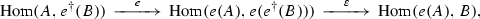and to prove the proposition, we need to check that this map is an isomorphism. To do this, evaluate $${\text {Hom}}(A,e^\dagger (B))$$ by the spectral sequence of Corollary 8.11. Its $$E_1$$-term is described in Proposition 9.7, and it coincides with the standard cobar-complex for the comonad $$R^\cdot \Phi$$ on the additive category $${\text {Ho}}(\mathcal {C})$$. Therefore the higher homology groups $$H^n(\Delta P,{\mathcal {H}{} { om}}(A,e^\dagger (B))$$, $$n \ge 1$$ vanish, so that the spectral sequence collapses at $$E_2$$, and then by Lemma 5.13, the homology in degree 0 is exactly $${\text {Hom}}(\mathsf{ev}(A),\overline{F}_\natural (\mathsf{ev}(B)) = {\text {Hom}}(e(A),B)$$. $$\square$$

### A model for spectra

As an application of the technology of stable comonads, let us sketch a simple model for the stable homotopy category based on chain complexes.

Denote by $${\text {Ab}}$$ the category of abelian groups. Recall that the Dold–Kan construction provides a pair of inverse equivalences

\begin{aligned} N:\Delta ^o {\text {Ab}}\rightarrow C_{\ge 0}({\text {Ab}}), \qquad D:C_{\ge 0}({\text {Ab}}) \rightarrow \Delta ^o{\text {Ab}}\end{aligned}
(10.3)

between the category $$\Delta ^o{\text {Ab}}$$ of simplicial abelian groups and the category $$C_{\ge 0}({\text {Ab}})$$ of chain complexes of abelian groups concentrated in non-negative homological degrees. For any functor $$F:{\text {Ab}}\rightarrow {\text {Ab}}$$, one can then define a functor

\begin{aligned} F_\cdot = N \circ F_{\Delta ^o} \circ D:C_{\ge 0}({\text {Ab}}) \rightarrow C_{\ge 0}({\text {Ab}}), \end{aligned}

where $$F_{\Delta ^o}$$ stands for the functor F applied pointwise to a simplicial abelian group. It is known that the functor $$F_\cdot$$ sends chain-homotopic maps to chain-homotopic maps, and for any complex $$E_\cdot \in C_{\ge 0}({\text {Ab}})$$, we have a functorial map

\begin{aligned} F_\cdot (E_\cdot ) \rightarrow F_\cdot (E_\cdot ). \end{aligned}
(10.4)

Moreover, if for some $$n \ge 0$$, a chain complex $$E_\cdot$$ is trivial in homological degrees $$< n$$ (that is, $$E_m = 0$$ for $$m < n$$), then $$F_\cdot (E_\cdot )$$ is also trivial in homological degree $$<n$$. Therefore one can define the stabilization $${\text {Stab}}(F)_\cdot$$ of $$F_\cdot$$ by setting

\begin{aligned} {\text {Stab}}(F)_\cdot (E_\cdot ) = \mathsf{lim}_{\overset{n \ge 0}{\rightarrow }}F_\cdot (E_\cdot [n])[-n], \end{aligned}
(10.5)

where the limit is taken with respect to the maps (10.4). By definition, the functor $${\text {Stab}}(F)_\cdot$$ commutes with homological shifts. Therefore we can extend it further to an endofunction of the category $$C_\cdot ({\text {Ab}}) \supset C_{\ge 0}({\text {Ab}})$$ of all chain complexes of abelian groups by setting

\begin{aligned} {\text {Stab}}(F)_\cdot (E_\cdot ) = \mathsf{lim}_{\overset{n \ge 0}{\rightarrow }}{\text {Stab}}(\tau _{\le -n}(E_\cdot )[n])[-n], \end{aligned}
(10.6)

where for any integer n, $$\tau _{\le n}E_\cdot$$ is the canonical truncation of the complex $$E_\cdot$$ at the homological degree n. Moreover, the stablization construction is compatible with compositions, in that for any two functors $$F,F':{\text {Ab}}\rightarrow {\text {Ab}}$$ that commute with filtered colimits, we have $${\text {Stab}}(F \circ F')_\cdot \rightarrow {\text {Stab}}(F)_\cdot \circ {\text {Stab}}(F')_\cdot$$, and this isomorphism is associative and unital in the obvious sense. In particular, if F is a comonad on $${\text {Ab}}$$, then $${\text {Stab}}(F)_\cdot$$ is a comonad on $$C_\cdot ({\text {Ab}})$$.

Now denote by $${\text {Sets}}_+$$ the category of pointed sets, and consider the forgetful functor $${\text {Ab}}\rightarrow {\text {Sets}}_+$$ sending an abelian group E to its underlying set with distinguished element $$0 \in E$$. This functor has a left-adjoint sending a pointed set $$\langle X,o \rangle$$ to the quotient $$\mathsf{Span}(X) = {\mathbb {Z}}[X]/{\mathbb {Z}}\cdot o$$ of the free abelian group $${\mathbb {Z}}[X]$$ generated by X by the subgroup spanned by the distinguished element $$o \in X$$. Thus $$E \rightarrow \mathsf{Span}(E)$$ is a comonad on $${\text {Ab}}$$, and this comonad obviously commutes with filtered colimits. Denote by $$Q = {\text {Stab}}(\mathsf{Span})$$ the corresponding comonad on $$C_\cdot ({\text {Ab}})$$, and equip $$C_\cdot ({\text {Ab}})$$ with the projective model structure of Example 2.13, so that $$\langle C_\cdot ({\text {Ab}}),C_\cdot ({\text {Ab}}) \rangle$$ is a stable model pair.

### Lemma 10.4

The comonad Q on $$\langle C_\cdot ({\text {Ab}}),C_\cdot ({\text {Ab}}) \rangle$$ is stable in the sense of Definition 10.2.

### Proof

By definition, Q commutes with filtered colimits. On one hand, a filtered colimit of quasiisomorphisms resp. fibrations in $$C_\cdot ({\text {Ab}})$$ is a quasiisomorphism resp. fibration, and on the other hand, any fibration resp. trivial fibration f in $$C_\cdot ({\text {Ab}})$$ can be represented as a filtered colimit of fibrations resp. trivial fibrations $$f_n:E_\cdot \rightarrow E_\cdot '$$ such that $$E_m=E_m'=0$$ for $$m \gg 0$$. Thus it suffices to prove that for any n, Q is a stable comonad on the full subcategory $$C_{\le n}({\text {Ab}}) \subset C_\cdot ({\text {Ab}})$$ of complexes $$E_\cdot$$ with $$E_m=0$$ for $$m \ge n$$. Since Q commutes with homological shifts, it actually suffices to consider the case $$n=0$$, when $${\text {Stab}}$$ is given by (10.5). Then the fact that Q sends fibrations to fibrations immediately follows from the fact that $$\mathsf{Span}$$ sends surjective maps to surjective maps, and the fact that Q is stable and sends quasiisomorphisms to quasiisomorphisms is well-known. $$\square$$

As a corollary of Lemma 10.4, we have the triangulated category of derived coalgebras $${\text {DCoalg}}(C_\cdot ({\text {Ab}}),Q)$$ of Definition 10.2, with the conservative forgetful functor $$e:{\text {DCoalg}}(C_\cdot ({\text {Ab}}),Q) \rightarrow {\mathcal {D}}({\text {Ab}})$$ to the derived category $${\mathcal {D}}({\text {Ab}}) \cong {\text {Ho}}(C_\cdot ({\text {Ab}}))$$ of the category of abelian groups. Proposition 10.3 then provides the right-adjoint functor $$e^\dagger :{\mathcal {D}}({\text {Ab}}) \rightarrow {\text {DCoalg}}(C_\cdot ({\text {Ab}}),Q)$$. Since $$C_\cdot ({\text {Ab}})$$ has arbitrary sums, so does the category $${\text {DCoalg}}(C_\cdot ({\text {Ab}}),Q)$$. Moreover, consider the standard t-structure on $${\mathcal {D}}({\text {Ab}})$$, and for any integer n, denote by $${\text {DCoalg}}^{\le n}(C_\cdot ({\text {Ab}}),Q) \subset {\text {DCoalg}}(C_\cdot ({\text {Ab}}),Q)$$ the full subcategory spanned by objects E such that e(E) lies in $${\mathcal {D}}^{\le n}({\text {Ab}})$$ (that is, $$e(E) \cong \tau _{\le n}e(E)$$). Let

\begin{aligned} {\text {DCoalg}}^-(C_\cdot ({\text {Ab}}),Q) = \bigcup _n {\text {DCoalg}}^{\le n}(C_\cdot ({\text {Ab}}),Q) \subset {\text {DCoalg}}(C_\cdot ({\text {Ab}}),Q) \end{aligned}

be the union of these subcategories (or equivalently, the full subcategory spanned by E such that $$e(E) \in {\mathcal {D}}({\text {Ab}})$$ is cohomologically bounded from above).

### Lemma 10.5

1. (i)

Assume given an object $$S \in {\text {DCoalg}}(C_\cdot ({\text {Ab}}),Q)$$ such that $$e(S) = {\mathbb {Z}}\in {\text {Ab}}\subset {\mathcal {D}}({\text {Ab}})$$. Then $${\text {Hom}}(S,E)=0$$ for any $$E \in {\text {DCoalg}}^{\le -1}(C_\cdot ({\text {Ab}}),Q)$$.

2. (ii)

The subcategories $${\text {DCoalg}}^{\le n}(C_\cdot ({\text {Ab}}),Q)$$, $$n \in {\mathbb {Z}}$$ form a non-degenerate t-structure on $${\text {DCoalg}}^-(C_\cdot ({\text {Ab}}),Q)$$. The forgetful functor e and its adjoint functor $$e^\dagger$$ are right t-exact. The functor e induces an equivalence between the heart of the t-structure on $${\text {DCoalg}}^-(C_\cdot ({\text {Ab}}),Q)$$ and the heart $${\text {Ab}}= {\mathcal {D}}^{\le 0}({\text {Ab}}) \cap {\mathcal {D}}^{\ge 0}({\text {Ab}}) \subset {\mathcal {D}}({\text {Ab}})$$ of the standard t-structure on $${\mathcal {D}}({\text {Ab}})$$.

### Proof

For (i), note that for any complex $$E_\cdot \in C_\cdot ({\text {Ab}})$$, the counit map $$Q(E_\cdot ) \rightarrow E_\cdot$$ is surjective, and let $$\overline{Q}(E_\cdot )$$ be its kernel. Then $$\overline{Q}$$ is a non-unital comonad on $$C_\cdot ({\text {Ab}})$$, it preserves quasiisomorphisms, and it is a well-known property of the functor Q that $$\overline{Q}$$ sends $${\mathcal {D}}^{\le n}({\text {Ab}})$$ into $${\mathcal {D}}^{\le n-1}({\text {Ab}})$$ for any integer n. Then to compute $${\text {Hom}}(S,E)$$, we can use the fundamental spectral sequence of Corollary 8.11. Its $$E_1$$-term is the standard cobar complex of the unital comonad Q, and it is quasiisomorphic to the cobar complex of the non-unital comonad $$\overline{Q}$$ with terms $${\text {Hom}}({\mathbb {Z}},\overline{Q}^n(e(E))[-n])$$. All these terms vanish for dimension reasons.

For (ii), note that the functor e is right t-exact by definition, and by construction, $$e^\dagger \circ e \cong {\text {Ho}}(Q)$$ is also right t-exact; since e is conservative, this means that $$e^\dagger$$ is right t-exact. By induction, to prove that the categories $${\text {DCoalg}}^{\le n}(C_\cdot ({\text {Ab}}),Q)$$ form a non-degenerate t-structure, it suffices to prove that for any integer n, the embedding $${\text {DCoalg}}^{\le n-1}(C_\cdot ({\text {Ab}}),Q) \subset {\text {DCoalg}}^{\le n}(C_\cdot ({\text {Ab}}),Q)$$ admits a right-adjoint. This is stable under shifts, so it suffices to take $$n=0$$. Then for any $$E \in {\text {DCoalg}}^{\le 0}(C_\cdot ({\text {Ab}}),Q)$$, the object $$e^\dagger (\tau _{\ge 0}e(E))$$ is right-orthogonal to $${\text {DCoalg}}^{\le -1}(C_\cdot (A\mathsf{B}),Q)$$ by adjunction, so it suffices to prove that the cone of the adjunction map $$E \rightarrow e^\dagger (\tau _{\ge 0}e(E))$$ lies in $${\text {DCoalg}}^{\le -1}(C_\cdot ({\text {Ab}}),Q)$$. This, together with the claim about the hearts, amounts to checking that for any $$E \in {\mathcal {D}}^{\le 0}({\text {Ab}})$$, the adjunction map $$Q(M) = e(e^\dagger (E)) \rightarrow E$$ is an isomorphism in homological degree 0. This is equivalent to saying that $$\overline{Q}$$ sends $${\mathcal {D}}^{\le 0}({\text {Ab}})$$ into $${\mathcal {D}}^{\le -1}({\text {Ab}})$$. $$\square$$

### Comparison

Now let us show how that category $${\mathcal {D}}(\mathcal {C}_\cdot ({\text {Ab}}),Q)$$ is related to spectra. Recall that a spectrum is what represents a generalized cohomology theory, and in the most classic model, it is given by a collection $$\{X_\cdot \}$$ of pointed simplicial sets $$X_n$$, $$n \ge 0$$ equipped with maps $$\delta _n:\Sigma X_n \rightarrow X_{n+1}$$, where $$\Sigma X = S^1 \wedge X$$ is the suspension functor (smash-product with the standard simplicial circle $$S^1$$). A map between two such collections $$\{X_\cdot \}$$, $$\{Y_\cdot \}$$ is a collection of maps $$X_n \rightarrow Y_n$$ that commute with the maps $$\delta _n$$, a map is a weak equivalence iff all the component maps $$X_n \rightarrow Y_n$$ are weak equivalences, and inverting weak equivalences via any of the many model structures present in the literature, one obtains the triangulated stable homotopy category $$\mathsf{StHom}$$.

The sphere spectrum S is formed by the spheres $$S^n$$ with the tautological maps $$\delta _n$$. For any spectrum X, its homotopy groups are given by $$\pi _n(X) = {\text {Hom}}(S,X[-n])$$, and a spectrum is $$X=\{X_\cdot \}$$ is connective if $$\pi _n(X)=0$$ for $$n < 0$$. Denote by $$\mathsf{StHom}^{\le 0} \subset \mathsf{StHom}$$ the full subcategory spanned by connective spectra. Then the embedding $$\mathsf{StHom}^{\le 0} \rightarrow \mathsf{StHom}$$ admits a right-adjoint functor sending a spectrum to its connective cover, so that the subcategories $$\mathsf{StHom}^{\le n} = \mathsf{StHom}^{\le 0}[-n] \subset \mathsf{StHom}$$, $$n \in {\mathbb {Z}}$$ form a t-structure on $$\mathsf{StHom}$$. The t-structure is not non-degenerate: while the intersection of all the $$\mathsf{StHom}^n \subset \mathsf{StHom}$$, $$n \in {\mathbb {Z}}$$ is trivial, their union

\begin{aligned} \mathsf{StHom}^- = \bigcup _m \mathsf{StHom}^{\le n} \subset \mathsf{StHom}\end{aligned}

is strictly smaller that $$\mathsf{StHom}$$. A spectrum is bounded below if it lies in $$\mathsf{StHom}^- \subset \mathsf{StHom}$$.

The category $$\mathsf{StHom}$$ has arbitrary sums, and it has the following remarkable property: for any triangulated category $${\mathcal {D}}$$ with arbitrary sums, a triangulated functor $$\nu :\mathsf{StHom}\rightarrow {\mathcal {D}}$$ that commutes with arbitrary sums admits a right-adjoint $$\nu ^\dagger$$ (this is a part of Brown Representability Theorem).

Further, recall that the reduced chain complex $$C_\cdot (X)$$ of a pointed simplicial set $$X \in \Delta ^o{\text {Sets}}_+$$ is given by

\begin{aligned} C_\cdot (X) = N\left( \mathsf{Span}_{\Delta ^o}(X)\right) , \end{aligned}

where N is the Dold-kand equivalence (10.3). We have the natural Künneth quasiisomorphism $$C_\cdot (X) \cong C_\cdot (\Sigma X)$$, and for any spectrum $$\{X_\cdot \}$$, we can define its homology complex $$C_\cdot (X_\cdot )$$ as

\begin{aligned} C_\cdot (X_\cdot ) = \mathsf{lim}_{\overset{n}{\rightarrow }}C_\cdot (X_n)[-n], \end{aligned}
(10.7)

where the limit is taken with respect to the maps $$\delta _n$$. Passing to the homotopy categories, we obtain the (ordinary) homology functor

\begin{aligned} H:\mathsf{StHom}\rightarrow {\text {Ho}}(C_\cdot ({\text {Ab}})) = {\mathcal {D}}({\text {Ab}}) \end{aligned}
(10.8)

from $$\mathsf{StHom}$$ to the derived category $${\mathcal {D}}({\text {Ab}})$$ of the category of abelian groups. For example, for the sphere spectrum S, we have

\begin{aligned} H(S) \cong {\mathbb {Z}}\in {\text {Ab}}= {\mathcal {D}}^{\le 0}({\text {Ab}}) \cap {\mathcal {D}}^{\ge 0}({\text {Ab}}). \end{aligned}
(10.9)

In general, the functor (10.8) is triangulated, and it commutes with arbitrary sums, so it admits a right-adjoint functor $$H^\dagger$$ by Brown representability. One can describe $$H^\dagger$$ in terms of the Dold–Kan equivalence (10.3): for any complex $$E_\cdot \in C_\cdot ({\text {Ab}})$$, the spectrum $$H^\cdot (E_\cdot )$$ has terms

\begin{aligned} H^\dagger (E_\cdot )_n = D(\tau _{\le 0}E_\cdot [n]), \end{aligned}
(10.10)

with the structure maps $$\delta _n$$ given by the maps (10.4). The functor H is right t-exact. Moreover, by Hurewicz Theorem, a spectrum $$E \in \mathsf{StHom}^-$$ lies in $$\mathsf{StHom}^{\le 0}$$ if and only if H(E) lies in $${\mathcal {D}}^{\le 0}({\text {Ab}}) \subset {\text {Ab}}$$, H is conservative on $$\mathsf{StHom}^-$$, and it induces an equivalence between the heart of the t-structure on $$\mathsf{StHom}$$ and the category $${\text {Ab}}= {\mathcal {D}}^{\le 0}({\text {Ab}}) \cap {\mathcal {D}}^{\ge 0}({\text {Ab}}) \subset {\mathcal {D}}({\text {Ab}})$$.

Now note that by adjunction, for any pointed simplicial set X, the simplicial abelian group $$\mathsf{Span}_{\Delta ^o}(X)$$ is a coalgebra over the comonad $$\mathsf{Span}_{\Delta ^o}$$ on $$\Delta ^o{\text {Ab}}$$, so that we have a natural structure map $$\alpha :C_\cdot (X) \rightarrow \mathsf{Span}_\cdot (C_\cdot (X))$$, and composing this map with the natural map $$\mathsf{Span}_\cdot \rightarrow {\text {Stab}}_0(\mathsf{Span}_\cdot )$$, we turn $$C_\cdot (X)$$ into a coalgebra over the comonad Q. Since Q commutes with homological shifts, for every spectrum $$\{X_\cdot \}$$, the shifts $$C_\cdot (X_n)[-n]$$ are also coalgebras over Q, and the transition maps in (10.7) are coalgebra maps, so that $$C_\cdot (X_\cdot )$$ is also naturally a coalgebra over Q. For example, for any chain complex $$E_\cdot \in C_\cdot ({\text {Ab}})$$, we have a natural isomorphism

\begin{aligned} C_\cdot (H^\dagger (E_\cdot )) \cong Q(E_\cdot ), \end{aligned}
(10.11)

where $$H^\dagger (E_\cdot )$$ is represented by the spectrum (10.10). Since the homology functor (10.8) is triangulated, sending a spectrum $$\{X_\cdot \}$$ to the derived coalgebra over Q corresponding to ordinary coalgebra $$C_\cdot (X_\cdot )$$ provides a triangulated functor

\begin{aligned} h:\mathsf{StHom}\rightarrow {\text {DCoalg}}(C_\cdot ({\text {Ab}}),Q) \end{aligned}
(10.12)

to the triangulated category of Definition 10.2 corresponding to the stable comonad Q of Lemma 10.4.

### Theorem 10.6

The functor (10.12) induces an equivalence of categories between $$\mathsf{StHom}^- \subset \mathsf{StHom}$$ and $${\text {DCoalg}}^-(C_\cdot ({\text {Ab}}),Q) \subset {\text {DCoalg}}(C_\cdot ({\text {Ab}}),Q)$$.

### Proof

By definition, we have $$H \cong e \circ h$$, where $$e:{\text {DCoalg}}(C_\cdot ({\text {Ab}}),Q) \rightarrow {\mathcal {D}}({\text {Ab}})$$ is the forgetful functor. The functor e has a right-adjoint functor $$e^\dagger$$, thus commutes with arbitrary sums, and then since e is conservative, this implies that h also commutes with arbitrary sums. Therefore by Brown representability, it has a right-adjoint functor $$h^\dagger$$, and we have $$H^\dagger \cong h^\dagger \circ e^\dagger$$.

Now, it immediately follows from (10.10) that the natural map $$H \circ H^\dagger \rightarrow e \circ e^\dagger$$ induced by the adjunction map $$h \circ h^\dagger \rightarrow \mathsf{Id}$$ is an isomorphism. Therefore h and $$h^\dagger$$ induce inverse equivalences between the smallest triangulated subcategories in $$\mathsf{StHom}$$ resp. $${\text {DCoalg}}(C_\cdot ({\text {Ab}}),Q)$$ that contain the images of the functors $$H^\dagger$$ resp. $$e^\dagger$$. Moreover, h and $$h^\dagger$$ induce equivalences between the hearts of the t-structures on $$\mathsf{StHom}^-$$ and $${\text {DCoalg}}^-(C_\cdot ({\text {Ab}}),Q)$$, and by induction, they also give equivalences

\begin{aligned}&\mathsf{StHom}^{\le n} \cap \mathsf{StHom}^{\ge m}\nonumber \\&\quad \cong {\text {DCoalg}}^{\le n}(C_\cdot ({\text {Ab}}),Q) \cap {\text {DCoalg}}^{\ge m}(C_\cdot ({\text {Ab}}),Q) \end{aligned}
(10.13)

for any integers $$n \ge m$$. Furthermore, since e and H are right t-exact and e is conservative, h is also right t-exact. By virtue of (10.13), this implies that for any integers $$m < n$$ and any $$E \in \mathsf{StHom}^{\le n}$$, $$h(\tau _{\ge m}(E)) \cong \tau _{\ge m}(h(E))$$. On the other hand, by Lemma 10.5 (i) and (10.9), $$h^\dagger$$ is also right t-exact, and (10.13) implies that $$\tau _{\ge m}h^\dagger (E) \cong h^\dagger (\tau _{\ge m}E)$$ for any $$E \in {\text {DCoalg}}(C_\cdot ({\text {Ab}}),Q)$$. Thus for any $$E \in \mathsf{StHom}^{\le n}$$, the adjunction map $$E \rightarrow h^\dagger (h(E))$$ reduces to the adjunction map for $$\tau _{\ge m}E$$ after applying $$\tau _{\ge m}$$, thus becomes invertible by (10.13), and since m is arbitrary, it must be invertible to begin with. Analogously, the adjunction map $$h(h^\dagger (E)) \rightarrow E$$ is invertible for any $$E \in {\text {DCoalg}}^{\le n}(C_\cdot ({\text {Ab}}),Q)$$, so that h and $$h^\dagger$$ are mutually inverse equivalences between $$\mathsf{StHom}^{\le n}$$ and $${\text {DCoalg}}^{\le n}(C_\cdot ({\text {Ab}}),Q)$$. Since n is arbitrary, the same holds for $$\mathsf{StHom}^-$$ and $${\text {DCoalg}}^-(C_\cdot ({\text {Ab}}),Q)$$. $$\square$$

### Remark 10.7

Since the category $${\text {DCoalg}}(C_\cdot ({\text {Ab}}),Q)$$ comes from a stable model pair, it has a spectral enrichment of Lemma 9.8. It is not difficult to show that it is compatible with the equivalence (10.12); we do not do it to save space.

### Remark 10.8

Theorem 10.6 is of course only useful if one can get good control over the functor Q, and it looks like it is much too big for that. Thus we mostly include Theorem 10.6 as a proof-of-concept and an illustration for Definition 10.2. We expect derived coalgebras to be much more useful when comonads in questions are polynomial functors (such as the cyclic power functor considered in ).

## DG categories

### Linear structures

Fix a commutative ring k, and denote by $$C_\cdot (k)$$ the unital symmetric monoidal category of complexes of k-modules. Recall (see e.g. ) that a DG category over k is a category $$A_\cdot$$ enriched over $$C_\cdot (k)$$ (that is, for any two objects $$a,a' \in A_\cdot$$, we have a complex of morphisms $$A_\cdot (a,a')$$). The category $$C_\cdot (k)$$ itself is a DG category in a natural way. A module over $$A_\cdot$$ is a functor $$F:A_\cdot ^o \rightarrow C_\cdot (k)$$ enriched over $$C_\cdot (k)$$ (that is, we have a complex $$F(a) \in C_\cdot (k)$$ for any $$a \in A_\cdot$$ and a map of complexes $$A_\cdot (a,a') \rightarrow {\text {Hom}}^\cdot (F(a),F(a'))$$ for any $$a,a' \in A_\cdot$$, subject to the usual conditions). For any module $$M_\cdot$$ over $$A_\cdot$$ and any complex $$P_\cdot \in C_\cdot (k)$$, we have a natural module $$P_\cdot \otimes M_\cdot$$ given by

\begin{aligned} (P_\cdot \otimes M_\cdot )(a) = P_\cdot (a) \otimes _k M_\cdot (a), \qquad a \in A_\cdot . \end{aligned}
(11.1)

Denote by $$I \in C_\cdot (k)$$ the complex $$k \overset{\mathsf{id}}{\longrightarrow } k$$ placed in homological degrees 0 and 1, and note that we have a natural short exact sequence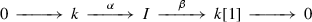(11.2)

in $$C_\cdot (k)$$. For any two modules $$M_\cdot$$, $$N_\cdot$$ over $$A_\cdot$$ and a map $$f:M_\cdot \rightarrow N_\cdot$$, the cone $${\text {Cone}}(f)_\cdot$$ is defined by taking the cartesian square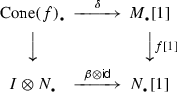(11.3)

pointwise over $$A_\cdot$$. By Yoneda, an object $$a \in A_\cdot$$ defines a module $$\mathsf{Y}(a)$$, $$\mathsf{Y}(a)(-) = A_\cdot (a',-)$$, and for any two objects $$a,a' \in A_\cdot$$, maps $$f:\mathsf{Y}(a) \rightarrow \mathsf{Y}(a')$$ are in a natural one-to-one correspondence with elements in the space $$Z_0(A_\cdot (a,a'))$$ of degree-0 cycles of the complex $$A_\cdot (a,a')$$.

For any DG category $$A_\cdot$$, one defines a category $$H_0(A_\cdot )$$ with the same objects, and homology classes $$f \in H_0(A_\cdot (-,-))$$ as morphisms. A DG category $$A_\cdot$$ is pre-triangulated if for any $$a,a' \in A_\cdot$$ and $$f \in Z^0(A_\cdot (a,a'))$$, $${\text {Cone}}(f)_\cdot \cong \mathsf{Y}(a'')$$ for some $$a'' \in A_\cdot$$. In this case, the category $$H_0(A_\cdot )$$ is canonically triangulated, with the shift defined by $$Y(a) = Y(a)$$, and distinguished triangles isomorphic to the triangles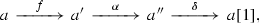where $$Y(a'') = {\text {Cone}}(f)_\cdot$$, $$\alpha$$ is induced by (11.2), and $$\delta$$ is induced by (11.3)

If a DG category $$A_\cdot$$ is small, then modules over $$A_\cdot$$ form a category; denote it by $$C_\cdot (A_\cdot )$$. The category $$C_\cdot (A_\cdot )$$ has a natural projective model structure whose weak equivalences W resp. fibrations F are pointwise quasiisomorphisms resp. pointwise degreewise surjections. Denote by $$C^{pf}_\cdot (A_\cdot ) \subset C_\cdot (A_\cdot )$$ the smallest full subcategory closed under weak equivalences, shifts and cones, and containing all the modules $$\mathsf{Y}(a)$$, $$a \in A_\cdot$$. It is elementary to check that $$C_\cdot (A_\cdot )$$ is a stable model category, and $$\langle C^{pf}_\cdot (A_\cdot ),C_\cdot (A_\cdot ) \rangle$$ is a stable model pair in the sense of Definition 2.12. The triangulated category $${\text {Ho}}(C_\cdot (A_\cdot ),W)$$ is denoted by $${\mathcal {D}}(A_\cdot )$$ and called the derived category of $$A_\cdot$$-modules, and $${\mathcal {D}}^{pf}(A_\cdot ) = {\text {Ho}}(C^{pf}_\cdot (A_\cdot )) \subset {\mathcal {D}}(A_\cdot )$$ is a full triangulated subcategory whose Karoubi envelope is the subcategory of compact objects in $${\mathcal {D}}(A_\cdot )$$. If $$A_\cdot$$ is pretriangulated, then the Yoneda functor $$a \mapsto \mathsf{Y}(a)$$ induces a triangulated equivalence $$H_0(A_\cdot ) \cong {\mathcal {D}}^{pf}(A_\cdot )$$.

More generally, if a pretriangulated DG category $$A_\cdot$$ is not small, it often happens that it contains a small full subcategory $$A'_\cdot$$ such that the Yoneda functor $$H^0(A_\cdot ) \rightarrow {\mathcal {D}}(A'_\cdot )$$, $$a \mapsto \mathsf{Y}(a)|_{A'_\cdot }$$ is fully faithful. In this case, $$H_0(A_\cdot )$$ also corresponds to a stable model pair—the one formed by $$C_\cdot (A'_\cdot )$$ and its full subcategory spanned by objects weakly equivalent to $$\mathsf{Y}(a)$$, $$a \in A_\cdot$$.

Conversely, assume given a stable model pair $$\langle \mathcal {C},\mathcal {C}' \rangle$$. Fix a commutative ring k, and denote by $$C^{pf}_\cdot (k) \subset C_\cdot (k)$$ the full subcategory spanned by finite-length complexes of finitely generated free k-modules. Note that $$C^{pf}_\cdot (k) \subset C_\cdot (k)$$ is a monoidal subcategory closed under finite coproducts, with the unit object k placed in degree 0. Moreover, $$C_\cdot (k)$$ carries the projective model structure, and all objects $$M_\cdot \in C^{pf}_\cdot (k) \subset C_\cdot (k)$$ are fibrant and cofibrant with respect to this model structure.

### Definition 11.1

A k -linear structure on $$\langle \mathcal {C},\mathcal {C}' \rangle$$ is a functor $$\otimes :C^{pf}_\cdot (k) \times \mathcal {C}' \rightarrow \mathcal {C}'$$ equipped with associativity isomorphisms

\begin{aligned} (M_\cdot \otimes N_\cdot ) \otimes X \cong M_\cdot \otimes (N_\cdot \otimes X), \qquad M_\cdot ,N_\cdot \in C^{pf}_\cdot (k),X \in \mathcal {C}\end{aligned}

and unitality isomorphisms $$k \otimes X \cong X$$, $$X \in \mathcal {C}$$, subject to the usual contraints, such that

1. (i)

for any $$M_\cdot$$, the functor $$M_\cdot \otimes -:\mathcal {C}' \rightarrow \mathcal {C}'$$ sends $$\mathcal {C}\subset \mathcal {C}'$$ into $$\mathcal {C}\subset \mathcal {C}'$$ and preserves finite limits and classes C, F, W, and

2. (ii)

for any fibrant and cofibrant $$X \in \mathcal {C}$$, the functor $$- \otimes X:C^{pf}_\cdot (k) \rightarrow \mathcal {C}\subset \mathcal {C}'$$ preserves finite products and classes C, F, W.

### Example 11.2

For any small DG category $$A_\cdot$$ over k, pointwise tensor product gives a canonical k-linear structure on $$\langle C^{pf}_\cdot (A_\cdot ),C_\cdot (A_\cdot ) \rangle$$.

Let now $$\langle \mathcal {C},\mathcal {C}' \rangle$$ be a stable model pair equipped with a k-linear structure, and define a DG category $$\mathcal {C}_\cdot$$ over k as follows:

1. (i)

objects of $$\mathcal {C}_\cdot$$ are fibrant and cofibrant objects $$X \in \mathcal {C}\subset \mathcal {C}'$$,

2. (ii)

for any two objects $$X,Y \in \mathcal {C}_\cdot$$, the complex $$\mathcal {C}_\cdot (c,c')$$ is given by

\begin{aligned} \mathcal {C}_i(X,Y) = \mathcal {C}(X,I[-i] \otimes Y), \qquad i \in {\mathbb {Z}}, \end{aligned}

where I is as in (11.2), the commutative ring k acts via its action on I, and the differential $$d:\mathcal {C}_{i+1}(X,Y) \rightarrow \mathcal {C}_i(X,Y)$$ is induced by the natural map $$\alpha [-(i+1)] \circ \beta [-i]:I[-i] \rightarrow I[-(i+1)]$$.

### Proposition 11.3

The DG category $$\mathcal {C}_\cdot$$ is pretriangulated, and the triangulated category $$H_0(\mathcal {C}_\cdot )$$ is naturally equivalent to $${\text {Ho}}(\mathcal {C},W)$$ as a triangulated category.

### Proof

For the first claim, note for any map $$f:X \rightarrow Y$$ in $$\mathcal {C}$$, we can take the cartesian square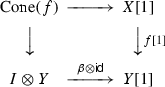(11.4)

in $$\mathcal {C}'$$, where we set $$X = k \otimes X$$, $$Y = k \otimes Y$$, and since the bottom map is a fibration by Definition 11.1 (ii), the square is also homotopy cocartesian. Thus $${\text {Cone}}(f)$$ lies in $$\mathcal {C}\subset \mathcal {C}'$$. If X and Y are fibrant and cofibrant, then so is $$I \otimes Y$$, hence also $${\text {Cone}}(f)$$, and by definition, we have $$Y({\text {Cone}}(f)) \cong {\text {Cone}}(\mathsf{Y}(f))$$.

For the second claim, recall that all objects $${\text {Ho}}(\mathcal {C},W)$$ can be represented by fibrant cofibrant objects in $$\mathcal {C}$$. Any map $$f:X \rightarrow Y$$ between two such objects by definition gives an element $$\widetilde{f} = \alpha \circ f \in \mathcal {C}_0(X,Y)$$, and since $$\beta \circ \alpha =0$$, $$\widetilde{f}$$ actually lies in $$Z_0(\mathcal {C}_\cdot (X,Y))$$. Moreover, let $$P = {\text {Cone}}(\beta [-1]) \cong k \oplus I[-1]$$, so that we have a decomposition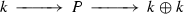(11.5)

of the form (2.3) of the diagonal map $$k \rightarrow k \oplus k$$. Then for two maps $$f_1,f_2:X \rightarrow Y$$, the cocycles $$\widetilde{f}_1,\widetilde{f}_2 \in Z_0(\mathcal {C}_\cdot (X,Y))$$ differ by a coboundary if and only if $$f_1 \oplus f_2:X \rightarrow Y \times Y \cong (k \oplus k) \otimes Y$$ factors through $$P \otimes Y$$. By Definition 11.1 (ii), tensoring (11.5) with Y gives a decomposition (2.3) for $$Y \rightarrow Y \times Y$$, so that this happens if and only if $$f_1$$ and $$f_2$$ represent the same map in $${\text {Ho}}(\mathcal {C},W)$$. This defines an equivalence $${\text {Ho}}(\mathcal {C},W) \cong H_0(\mathcal {C}_\cdot )$$.

Finally, to see that the equivalence is triangulated, recall that cones in $$H_0(\mathcal {C}_\cdot )$$ are defined by cartesian and homotopy cartesian squares (11.4), and these canonically extend to the fiber versions of diagrams (2.11). $$\square$$

### Gluing

It turns out that the notion of a k-linear structure is not strong enough for the gluing formalism of Sects. 3 and 9, and we have to modify it. Note that for any category $$\mathcal {C}$$ and small category I, a functor $$C_\cdot ^{pf}(k) \times \mathcal {C}\rightarrow \mathcal {C}$$ induces a functor $$C_\cdot ^{pf}(k) \times \mathcal {C}^I \times \mathcal {C}^I$$ for the functor category $$\mathcal {C}^I$$.

### Definition 11.4

A k-linear structure on a stable model pair $$\langle \mathcal {C},\mathcal {C}' \rangle$$ is left resp. right-continuous if the induced functor $$C_\cdot ^{pf}(k) \times \mathcal {C}^{} \rightarrow \mathcal {C}^{}$$ is a k-linear structure on the stable model pair $$\langle \mathcal {C}^{},\mathcal {C}^{'} \rangle$$, where $$\mathcal {C}^{'}$$ is equipped with the projective resp. injective model structure, and continuous if it is left and right-continuous.

Although it is not strictly necessary, it is also convenient to strengthen Definition 8.1.

### Definition 11.5

A k -linear stable model prefibration $$\langle \mathcal {C},\mathcal {C}' \rangle$$ over a category I is a stable model prefibration $$\langle \mathcal {C},\mathcal {C}' \rangle$$ over I in the sense of Definition 8.1 such that for any morphism $$f:i \rightarrow i'$$ in I, the transition functor $$f^*:\mathcal {C}'_{i'} \rightarrow \mathcal {C}_i$$ preserves finite limits and sends $$\mathcal {C}_{i'} \subset \mathcal {C}'_{i'}$$ into $$\mathcal {C}_i \subset \mathcal {C}'_i$$, equipped with a functor $$\otimes :C_\cdot ^{pf}(k) \times \mathcal {C}' \rightarrow \mathcal {C}'$$ cartesian over I, and associativity and unitality isomorphisms such that for any $$i \in \mathcal {C}_i$$, the induced functor $$\otimes :\mathcal {C}_\cdot ^{pf}(k) \times \mathcal {C}'_i \rightarrow \mathcal {C}'_i$$ is a continuous k-linear structure on $$\langle \mathcal {C}_i,\mathcal {C}'_i \rangle$$ in the sense of Definition 11.4.

We note that since $$f^*(\mathcal {C}_{i'}) \subset \mathcal {C}_i$$ for any $$f:i \rightarrow i'$$, the functor $$\mathcal {C}\rightarrow I$$ is also a prefibration, so that saying that $$\otimes$$ is cartesian over I makes sense. One probably could get away with weaker assumptions on $$f^*$$, but we do not know any interesting examples that would require larger generality. In particular, we do need the transition functors $$f^*$$ to commute with $$\otimes$$, and this already implies that they commute with finite sums and products. One immediate simplification that results from requiring the functors $$f^*$$ to preserve finite limits is that if I is a Reedy category and $$\langle \mathcal {C},\mathcal {C}' \rangle$$ is good in the sense of Definition 8.1, then $$\mathcal {C}'$$ satisfies the condition (i) of Theorem 7.17. Therefore there is no need to consider matching expansion—by Propositions 8.2 and 7.26, already $$\langle {\text {Sec}}(I,\mathcal {C}),{\text {Sec}}(I,\mathcal {C}') \rangle$$ is a stable model pair.

### Proposition 11.6

Assume given a stable model prefibration $$\langle \mathcal {C},\mathcal {C}' \rangle$$ over a Reedy category I that is good in the sense of Definition 8.1 and k-linear in the sense of Definition 11.5. Then the functor $$\otimes$$ induces a continuous k-linear structure in the sense of Definition 11.4 on the stable model pair $$\langle {\text {Sec}}(I,\mathcal {C}),{\text {Sec}}(I,\mathcal {C}') \rangle$$.

### Proof

Definition 11.1 (i) immediately follows from the definitions. To prove that $$\otimes$$ gives a k-linear structure, one has to check is Definition 11.1 (ii)—namely, the fact that $$\otimes$$ sends fibrations and cofibrations in $$C_\cdot ^{pf}(k)$$ to fibrations and cofibrations in $${\text {Sec}}(I,\mathcal {C})$$. The category $$C_\cdot ^{pf}(k)$$ is self-dual, and the duality interchanges fibrations and confibrations, so that it suffices to prove the statement for fibration. Moreover, by induction on degree and Proposition 3.12, it suffices to do it in the situation of Proposition 3.8, under the assumption that the functor $$\Phi$$ sends $$C_0 \subset \mathcal {C}_0'$$ into $$\mathcal {C}_1 \subset \mathcal {C}_1'$$ and preserves finite limits. Then an object $$\langle c_0,c_1,\alpha \rangle \in \mathsf{R}(\Phi )$$ is fibrant cofibrant iff $$c_0$$ is fibrant cofibrant in $$\mathcal {C}_0$$ and $$\alpha :c_1 \rightarrow \Phi (c_0)$$ is fibrant cofibrant in $$\mathcal {C}_1^{}$$, so that the claim immediately follows from Definition 11.1 (ii) for $$\mathcal {C}_0$$ and $$\mathcal {C}_1^{}$$. Thus $$\otimes$$ indeed gives a k-linear structure; to prove that it is continuous, apply the same argument to $$I \times $$. $$\square$$

Once we have fixed Definition 11.5, giving a k-linear version of stable model families of Definition 9.3 and stable comonads of Section 9.3 is completely straightforward. For a stable model family $$\langle \mathcal {C},\mathcal {C}' \rangle$$, we require that transition functor $$f_!$$ for a map $$f:i \rightarrow i'$$ sends $$\mathcal {C}_i$$ into $$\mathcal {C}_{i'}$$ and preserves finite limits, and that the functor $$\otimes$$ defining a k-linear structure is cocartesian over I. For a comonad $$\Phi$$ on a stable k-linear model pair $$\langle \mathcal {C},\mathcal {C}'\rangle$$, we require that $$\Phi (\mathcal {C}) \subset \mathcal {C}$$, $$\Phi$$ preserves finite limits, and we have an isomorphism $$\Phi \circ \otimes \cong \otimes \circ (\mathsf{Id}\times \Phi )$$ that is compatible with all the structure maps. Under these assumptions, Proposition 11.6 produces k-linear structures on the stable model pairs defining the categories $${\text {DRec}}(I,\mathcal {C})$$ resp. $${\text {DCoalg}}(\mathcal {C},\Phi )$$. All the general theory works in the k-linear case, too; in particular, we have the spectral sequence of Corollary 8.11.

### Remark 11.7

An example of a k-linear comonad on the category $$C_\cdot (k)$$ is given by $$\Phi (M_\cdot ) = {\text {Hom}}_k(A_\cdot , M_\cdot )$$, where $$A_\cdot$$ is a DG algebra termise-projective over k, and it is easy to see that under mild finiteness assumptions, all examples are of this form. In this case, coalgebras over $$\Phi$$ coincide with modules over $$A_\cdot$$, the fundamental spectral sequence of Corollary 8.11 reduces to the bar complex of the algebra $$A_\cdot$$, and derived coalgebras in our sense roughly correspond to $$A_\infty$$-modules. One can probably also incorporate $$A_\infty$$-algebras into the picture by considering weakly special k-linear prefibration over $$\Delta$$ but we did not go into this. Note that the fact that unitality requires special treatment and is best considered simply as a condition on the level of the derived category is well-known in the theory of $$A_\infty$$-algebras and $$A_\infty$$-modules (see e.g. , where the combinatorics is very close to our Lemma 10.1).

### Examples

Apart from DG categories, the main source of k-linear stable model pairs are k-linear abelian categories. If we have a k-linear abelian category $$\mathcal {E}$$ with enough projectives resp. injectives, then the category of chain complexes $$C_\cdot (\mathcal {E})$$ with the projective resp. injective model structure of Example 2.13 is a stable model category. For any abelian subcategory $$\mathcal {E}_0 \subset \mathcal {E}$$, we can consider the full subcategory $$C_\cdot (\mathcal {E},\mathcal {E}_0) \subset C_\cdot (\mathcal {E})$$ spanned by complexes with homology in $$\mathcal {E}_0$$, and then $$\langle C_\cdot (\mathcal {E},\mathcal {E}_0),C_\cdot (\mathcal {E}) \rangle$$ is a k-linear stable model pair. If we denote by $$C^{pf}_\cdot (\mathcal {E}) \subset C_\cdot (\mathcal {E})$$ the full subcategory spanned by perfect complexes, then $$\langle C_\cdot ^{pf}(\mathcal {E}),C_\cdot (\mathcal {E}) \rangle$$ is also a k-linear stable model pair.

For example, for any Noetherian scheme X / k, the category $${\text {QCoh}}(X)$$ of quasicoherent sheaves on X has enough injectives. If X is affine, the subcategory $${\text {Coh}}(X) \subset {\text {QCoh}}(X)$$ has enough projectives. In general, it does not, but it is still a full abelian subcategory in $${\text {QCoh}}(X)$$, so that we have a k-linear stable model pair $$\langle C_\cdot ({\text {QCoh}}(X),{\text {Coh}}(X)),C_\cdot ({\text {QCoh}}(X)) \rangle$$. The corresponding triangulated category is the derived category $${\mathcal {D}}_c(X)$$ of complexes with coherent homology, and it is in fact equivalent to the derived category $${\mathcal {D}}({\text {Coh}}(X))$$. Analogously, the k-linear stable model pair $$\langle C^{pf}_\cdot ({\text {QCoh}}(X)),C_\cdot ({\text {QCoh}}(X))\rangle$$ produces the derived category $${\mathcal {D}}^{pf}(X)$$ of perfect complexes on X.

The corresponding pretriangulated DG categories of Proposition 11.3 are quasiequivalent to the standard DG enhancements for $${\mathcal {D}}_c(X)$$ and $${\mathcal {D}}^{pf}(X)$$, and as far as categories are concerned, the theories seem completely parallel. However, they diverge when we start dealing with functors. Namely, consider the following situation.

### Example 11.8

Assume given two k-linear abelian categories $$\mathcal {E}_0$$, $$\mathcal {E}_1$$ with enough projectives resp. injectives, and a pair of k-linear functors $$e:\mathcal {E}_0 \rightarrow \mathcal {E}_1$$, $$e^\dagger :\mathcal {E}_1 \rightarrow \mathcal {E}_0$$ right-adjoint to e. Then by adjunction, e is right-exact and $$e^\dagger$$ is left-exact, and if we equip $$C_\cdot (\mathcal {E}_0)$$ resp. $$C_\cdot (\mathcal {E}_1)$$ with the projective resp. injective model structure, then termwise extensions of e and $$e^\dagger$$ are left resp. right-derivable and form a Quillen adjoint pair.

The situation of Example 11.8 occurs in geometry when one considers partial and categorical resolutions, such as e.g. . Namely, assume given an affine scheme Y / k and a proper map $$\pi :X \rightarrow Y$$. Then the pullback functor $$e=\pi ^*$$ and the pushforward functor $$e^\dagger =\pi _*$$ as as in Example 11.8. We now note that neither $$L^\cdot \pi ^*$$ nor $$R^\cdot \pi _*$$ has a natural enhancement to a DG functor, so that in the standard DG formalism, one can only consider these functors if one makes additional choices. In the stable model pair formalism, no choices are needed. What happens is, the DG category associated to a stable model pair in Proposition 11.3 consists of fibrant cofibrant objects, but while these span the homotopy category, they are not necessarily preserved by derived functors. Therefore not every stable left or right-derivable functor $$\Phi :\langle \mathcal {C},\mathcal {C}' \rangle \rightarrow \langle \mathcal {E},\mathcal {E}'\rangle$$ between k-linear stable model pairs descends to a DG functor $$\Phi _\cdot :\mathcal {C}_\cdot \rightarrow \mathcal {E}_\cdot$$ between the corresponding DG categories. In this respect, the formalism of stable model pairs is more flexible. Another example of this flexibility is the Fourier-Mukai transform of Example 3.5.

While functors of the form considered in Example 11.8 can be used in the elementary gluing construction of Proposition 3.8, their construction relies on using model structures of two different type on the source and the target, and this cannot be iterated. Here are two geometric examples of iterated gluing that use only one of the two model structures.

### Example 11.9

Assume given an affine simplicial scheme X over k. Then for any morphism $$f:[n'] \rightarrow [n]$$ in $$\Delta$$, we have the corresponding affine map $$X(f):X([n]) \rightarrow X([n'])$$, and the functor $$X(f)_*$$ is exact, hence right-derivable with respect to the projective model structures. Then the categories $$C_\cdot ({\text {QCoh}}(X([n])))$$ with the functors $$X(f)_*$$ form a k-linear stable model prefibration over $$\Delta$$ in the sense of Definition 11.5.

### Example 11.10

Assume given a small category I and a functor X from I to schemes. Then for any morphism $$f:i \rightarrow i'$$ in I, we have the map $$X(f):X(i) \rightarrow X(i')$$, and the functor $$X(f)_*$$ is right-derivable with respect to the injective model structures. The categories $$C_\cdot ({\text {QCoh}}(X(i)))$$ with the transition functors $$X(f)_*$$ form a k-linear stable model family over I.

In both Examples 11.9 and 11.10, one can of course restrict one’s attention to complexes of coherent sheaves, or perfect complexes, by considering the corresponding stable model pair (although one has to check that the transition functors preserve complexes in the chosen class). In Example 11.10, the category $${\text {DRec}}(I,C_\cdot ({\text {QCoh}}(X(i))))$$ corresponds pretty closely to the intuitive idea of the derived category of quasicoherent sheaves on a diagram of schemes. In the interests of full disclosure, we should mention that it is probably equivalent to the derived category $${\mathcal {D}}({\text {Rec}}(I,{\text {QCoh}}(X(i)))$$ of the abelian category $${\text {Rec}}(I,{\text {QCoh}}(X(i)))$$, although we did not check this.

For another series of examples, one can consider topological sheaves instead of coherent ones. For the simplest example, let X be a simplicial scheme over $$\mathbb {C}$$, and consider the categories $${\text {Shv}}(X([n])_{an},k)$$ of sheaves of k-modules on the underlying complex-analytic spaces $$X([n])_{an}$$. Then for any $$f:[n'] \rightarrow [n]$$, the pullback functor $$X(f)^*:{\text {Shv}}(X([n'])_{an},k) \rightarrow {\text {Shv}}(X([n])_{an},k)$$ is exact, thus right-derivable with respect to the injective model structure, and the categories $$C_\cdot ({\text {Shv}}(X([n])_{an},k))$$ with the transition functors $$X(f)^*$$ form a k-linear stable model prefibration over $$\Delta ^o$$. Again, one can restrict one’s attention to a subclass of sheaves by considering model pairs (for example, one can consider complexes of sheaves with constructible cohomology). The category $${\text {DSec}}(\Delta ^o,{\text {Shv}}(X_{an},k))$$ then gives a good notion of the category of complexes of sheaves on the simplicial scheme X. One natural example of a simplicial scheme is the stack quotient $$Y/\!/G$$ of a scheme Y by an action of an algebraic group G, understood as the simplicial scheme with terms $$X([n]) = Y \times G^n$$. In this case, $${\text {DSec}}(\Delta ^o,{\text {Shv}}(X_{an},k))$$ contains as a full subcategory the equivariant derived category $${\text {Shv}}_G(Y_{an},k)$$ of .

For étale sheaves with finite coefficients, exactly the same constructions work. A complex of étale sheaves with coefficients in $${\mathbb {Z}}_l$$ is usually defined as an inverse system of compatible complexes of $${\mathbb {Z}}/l^n{\mathbb {Z}}$$-sheaves, and this does not combine too well with injective model structures. However, one can equally well consider direct systems of complexes $$E^\cdot _n$$ over $${\mathbb {Z}}/l^n{\mathbb {Z}}$$ with maps $$E^\cdot _n \rightarrow E^\cdot _{n+1}$$ that induce quasiisomorphisms

\begin{aligned} E^\cdot _n \cong {\text {RHom}}^\cdot _{{\mathbb {Z}}/l^{n+1}{\mathbb {Z}}}\left( {\mathbb {Z}}/l^n{\mathbb {Z}},E^\cdot _{n+1}\right) , \qquad n \ge 1. \end{aligned}

Since $${\text {Hom}}(\mathbb {Q}_l/{\mathbb {Z}}_l,\mathbb {Q}_l/{\mathbb {Z}}_l)={\mathbb {Z}}_l$$, this gives the same derived category, but now the transition functors in the system are right-derivable, so that we have a $${\mathbb {Z}}_l$$-linear model family over the set of positive integers with the usual total order. Gluing this system can be done by the same general formalism, and then the resulting stable model pairs can be used for the geometric gluing situations such as sheaves on a simplicial scheme. There should also be parallel constructions for the categories of $${\mathcal {D}}$$-modules and cristals, but we did not pursue this.

## Localization

To finish the paper, let us construct the stable model pair counterpart of the Verdier quotient of Theorem 3.13. As we have remarked in Sect. 3.4, this is trivial in the semiorthogonal decomposition case. The simplest practically important example of a Verdier quotient that is not of this type is the following:

• $${\mathcal {D}}_0$$ is the bounded derived category of finite-dimensional vector spaces over a field k, and $${\mathcal {D}}\supset {\mathcal {D}}_0$$ is the derived category of all vector spaces (or at least, of vector spaces of countable dimension).

Since $${\mathcal {D}}_0$$ is small, it is localizing, so the quotient $${\mathcal {D}}/{\mathcal {D}}_0$$ is well-defined. It is known as the Calkin category of the field k, and its construction can be considerably generalized (for example, one can start with perfect modules over a arbitrary DG algebra $$A_\cdot$$, embed it into the category of all modules, and take the quotient). Another related class of examples is given by singular derived categories that appear, among other things, in the study of Tate cohomology and of matrix factorizations. Already in the simplest case, the Calkin category is quite useful—for example, it gives a short and clean approach to the Tate residue construction. It is definitely not of the semiorthogonal type, and in fact, the quotient functor $${\mathcal {D}}\rightarrow {\mathcal {D}}/{\mathcal {D}}_0$$ admits no sections at all (to see this, one can take an additive invariant in the sense of  such as K-theory or Hochschild homology, and observe that the connecting differential in the localization long exact sequence is non-trivial, and in fact an isomorphism). Keeping this example in mind, let us turn to stable model pairs.

### Generalities

Historically, the first localization construction in the model category setting was given by Bousfield  who wanted to avoid enlarging universes when localizing the stable homotopy category. While  only deals with spaces and spectra, the approach has been generalized to a large class of stable and unstable model categories, and this generalization is now known as “Bousfield localization”. Roughly speaking, one starts with a model category $$\mathcal {C}$$, enlarges the class W of weak equivalences just as in Theorem 3.13, keeps one of the two classes C or F, say C, defines F by the lifting property, and then uses a general machine to show that under certain assumptions, this gives a new model structure on $$\mathcal {C}$$. The most difficult part seems to be Definition 2.6 (iv), that is, the existence of fibrant replacements. These are obtained by transfinite induction using the so-called “small object argument”.

In principle, this could be done in our setting of stable model pairs, and due to the way we have formulated Definition 2.12, this is even simpler than the general case—no transfinite induction is necessary, and a fibrant replacement can be obtained in one step.

However, this does not solve our problem, since it does not cover all the applications that occur in real life. For example, a procedure of Bousfield type can never apply to the Calkin category example.

The problem is exactly fibrant replacements. Since one has enlarged W, hence also $$C \cap W$$, F has shrunk, and fibrant objects are no longer fibrant with respect to the new model structure. To correct for this, one replaces an object X with the filtered colimit over an appropriate category I of objects $$X'$$ equipped with a map $$f:X \rightarrow X'$$ in the class $$C \cap W$$. In the stable case, being in W means that the cone of f is in the subcategory $${\mathcal {D}}_0$$, and the I must be large enough to be cofinal after projecting to the category W(X) of (3.8). In the Calkin case, this means that I is infinite, and the colimit is not in W(X) anymore.

A moment’s reflection shows that the problem is not specific to the Calkin category. Indeed, by its very definition, the Bousfield localization functor is the derived functor of $$\mathsf{Id}:\mathcal {C}\rightarrow \mathcal {C}$$, and then by Quillen Adjunction Theorem, it comes equipped with an adjoint. In the stable situation, we are then back in the case of a semiorthogonal decomposition (and then if we work with stable pairs and not just stable model categories, the localization procedure is not needed at all since there is a simpler alternative).

If one treats this problem as a sign of deficiency in the axioms of a model category, then two possible solutions come to mind.

1. (I)

Definition 2.6 is too weak. One should simply impose whatever one wants for Bousfield localization, e.g. the existence of all limits, the fact that the category is “cofibrantly generated”, and so on.

2. (II)

Definition 2.6 is too strong. One could envision a notion of a pre-model structure on a saturated relative category $$\langle \mathcal {C},W \rangle$$ consisting of two classes of maps $$\overline{C}$$, $$\overline{F}$$ such that both are closed under compositions, $$W = \overline{F} \cdot \overline{C}$$, maps in $$\overline{F}$$ admit pullbacks and are closed under pullbacks, and maps in $$\overline{C}$$ admit pushouts and are closed under pushouts. This already allows one to show that maps in $${\text {Ho}}(\mathcal {C},W)$$ are reduced to diagrams (2.1) of length 3, and gives some control over the localization (in particular, one can impose a version of the stability condition and then prove that $${\text {Ho}}(\mathcal {C},W)$$ is triangulated).

Option (I) has become de facto standard in much of the literature, starting from  (where one even requires that factorizations in Definition 2.6 (iv) are functorial, with no justification given except for sheer laziness). It does not work for our purposes at all since it completely excludes several huge classes of applications. Option (II) is tempting. Basically, it axiomatizes the structure present on a category $$\mathcal {C}$$ equipped with a model embedding $$\mathcal {C}\subset \mathcal {C}'$$, with $$\overline{C}=C \cap W$$ and $$\overline{F} = F \cap W$$. To insure that $$\langle \mathcal {C},W \rangle$$ is localizable, it is probably prudent to impose a set-theoretical condition. For example, for any map g, one can consider the categories of factorizations $$g = g' \circ c$$, $$g = f \circ g'$$, $$c \in \overline{C}$$, $$f \in \overline{F}$$, and one could ask that they have cofinal small subcategories. Definition 2.6 (iv) would definitely insure it, but it seems like an overkill; so does requiring that $$\mathcal {C}$$ has all finite limits and colimits. Of course, in going this way, we lose the Quillen Adjunction Theorem, as we indeed must if we want to consider general Verdier quotients. Moreover, constructing derived functors would now involve taking certain filtered limits or colimits, and if the target category is too small, these need not exist. But this might just correspond to the nature of things—after all, the situation for abelian categories is exactly the same, see e.g. .

However, completely dispensing with model categories and developing a replacement such as (II) above from scratch would be a huge enterprise, and at present, we are not ready to do it.

So, in this paper, we adopt a third option. We keep the foundations exactly as they are, but we recall that we work with model pairs, and we use the freedom to enlarge the ambient category $$\mathcal {C}' \supset \mathcal {C}$$. To do this, we need the technology of inductive completions.

### Inductive completions

Assume given a category $$\mathcal {C}$$. Recall that inductive completion $$\mathsf{Ind}\,\mathcal {C}$$ of $$\mathcal {C}$$ is the category whose objects are pairs $$\langle I,X \rangle$$ of a filtered small category I and a functor $$X:I \rightarrow \mathcal {C}$$, with morphisms from $$\langle I,X \rangle$$ to $$\langle X',I' \rangle$$ given by

\begin{aligned} {\text {Hom}}(\langle I,X \rangle ,\langle I',X' \rangle ) = \mathsf{lim}_I\mathsf{colim}_{I'}{\text {Hom}}(X(i),X'(i')). \end{aligned}

The original reference for inductive completions is , and a good recent overview with complete proofs is [29, Chapter 6]. Let us recall some basic general facts.

For any $$\langle I,X \rangle \in \mathsf{Ind}\,\mathcal {C}$$ and cofinal functor $$\varphi :I' \rightarrow I$$ with filtered $$I'$$, the tautological map $$\langle I',\varphi ^*X \rangle \rightarrow \langle I,X \rangle$$ is an isomorphism. In particular, for every filtered small category $$I'$$, we have a cofinal functor $$I \rightarrow I'$$ from a category I such that $$I^o$$ is ordered in the sense of Definition 7.3 (for example, one can take the category $$I' = V(S)$$ of Example 7.2, where S is the set of objects in I). Therefore every object in $$\mathsf{Ind}\,\mathcal {C}$$ can be represented by a pair $$\langle I,X \rangle$$ with ordered $$I^o$$.

The category $$\mathsf{Ind}\,\mathcal {C}$$ has all filtered colimits. We have a natural embedding $$\mathcal {C}\rightarrow \mathsf{Ind}\,\mathcal {C}$$ sending $$c \in \mathcal {C}$$ to $$\langle \mathsf{pt},c \rangle$$, and this embedding is fully faithful. Any object $$c \in \mathcal {C}$$ is compact in $$\mathsf{Ind}\,\mathcal {C}$$ (that is, $${\text {Hom}}(c,-)$$ commutes with filtered colimits). Conversely, any compact $$\langle I,c \rangle \in \mathsf{Ind}\,\mathcal {C}$$ is a retract of an object c(i) for some $$i \in I$$. A functor $$F:\mathcal {C}\rightarrow \mathcal {C}'$$ defines a functor $$\mathsf{Ind}\,F:\mathsf{Ind}\,\mathcal {C}\rightarrow \mathsf{Ind}\,\mathcal {C}'$$ that restricts to F on $$\mathcal {C}\subset \mathsf{Ind}\,\mathcal {C}$$, and a morphism $$F \rightarrow F'$$ induces a morphism $$\mathsf{Ind}\,F \rightarrow \mathsf{Ind}\,F'$$. If $$\mathcal {C}$$ is small, $$\mathsf{Ind}\,\mathcal {C}$$ can be equivalently described as the smallest full subcategory in $${\text {Sets}}^{I^o}$$ containing the Yoneda image of $$\mathcal {C}$$ and closed under all filtered colimits. If $$\mathcal {C}$$ already has all filtered colimits, then we have a natural functor $$\mathsf{Ind}\,\mathcal {C}\rightarrow \mathcal {C}$$ sending $$\langle I,X \rangle$$ to $$\mathsf{colim}_I X$$. However, this functor is never an equivalence. In particular, “inductive completion” is abuse of terminology, since $$\mathsf{Ind}\,$$ is not an idempotent operation ( uses “indization”, we stick to the old term because it sounds better). For any small category I, we have an obvious functor

\begin{aligned} \mathsf{Ind}\,\mathcal {C}^I \rightarrow (\mathsf{Ind}\,\mathcal {C})^I, \end{aligned}
(12.1)

and if I is a finite ordered category, this functor is an equivalence of categories (this is [29, Theorem 6.4.3]). In particular, this holds for $$I = $$, and in this case, more is true: for any map $$f:\langle I,c \rangle \rightarrow \langle I',c' \rangle$$, there exists a filtered small category $$\widetilde{I}$$, two cofinal functors $$\pi :\widetilde{I} \rightarrow I$$, $$\pi ':\widetilde{I} \rightarrow \pi '$$, and a map $$\widetilde{f}:\pi ^*c \rightarrow \pi ^{'*}c'$$ such that $$\mathsf{colim}_{\widetilde{I}}\widetilde{f}=f$$ (this is [29, Proposition 6.1.13]).

### Lemma 12.1

If $$\mathcal {C}$$ has finite colimits, then $$\mathsf{Ind}\,\mathcal {C}$$ has all colimits. If $$\mathcal {C}$$ has finite limits, then $$\mathsf{Ind}\,\mathcal {C}$$ also has finite limits, and for any morphism $$f:\langle I',X' \rangle \rightarrow \langle I,X \rangle$$, the natural map

\begin{aligned} \mathsf{colim}_I(X_i \times _{\langle I,X \rangle } \langle I',X' \rangle ) \rightarrow \langle I',X' \rangle \end{aligned}
(12.2)

is an isomorphism.

### Proof

Since $$\mathsf{Ind}\,\mathcal {C}$$ has all filtered colimits, it suffices to check that it has colimits $$\mathsf{colim}_I$$ over a finite ordered category I. These exists in $$\mathcal {C}$$, so that we have an adjoint pair of functors $$\tau :\mathcal {C}\rightarrow \mathcal {C}^I$$, $$\mathsf{colim}_I:\mathcal {C}^I \rightarrow \mathcal {C}$$, with $$\tau$$ sending $$c \in \mathcal {C}$$ to the constant functor with value c. To extend this adjoint pair to $$\mathsf{Ind}\,\mathcal {C}$$ and $$(\mathsf{Ind}\,\mathcal {C})^I$$, it suffices to use the equivalence (12.1). For finite limits, the argument is exactly the same. Finally, to check that (12.2) is an isomorphism, note that f can be represented as colimit over $$I' \times I$$ of maps $$X_{i'} \times _{\langle I,X \rangle } X_i \rightarrow X_i$$, and we first take colimit over $$I'$$, we obtain exactly the map $$X_i \times _{\langle I,X \rangle } \langle I',X' \rangle \rightarrow X_i$$. $$\square$$

Now assume given a saturated relative category $$\langle \mathcal {C},W \rangle$$ with a model structure $$\langle C,F \rangle$$. Note that since  is a finite ordered category, every map in $$\mathsf{Ind}\,\mathcal {C}$$ can be represented by a filtered system $$\langle I,g \rangle$$ of maps in $$\mathcal {C}$$, and we can further assume that $$I^o$$ is ordered.

### Definition 12.2

A map in $$\mathsf{Ind}\,\mathcal {C}$$ lies in the class C, F, $$\overline{C}$$, $$\overline{F}$$, $$\overline{W}$$ iff it is a retract of a map given by a system $$\langle I,g \rangle$$ with ordered $$I^o$$ and g in the class C, F, $$C \cap W^I$$, $$F \cap W^I$$, $$W^I$$ with respect to the model structure of Lemma 7.3 on $$\langle \mathcal {C}^I,W^I \rangle$$. A map g in $$\mathsf{Ind}\,\mathcal {C}$$ lies in the class W if $$g=f \circ c$$ with $$f \in \overline{F}$$ and $$c \in \overline{C}$$.

### Lemma 12.3

Assume given a commutative square (2.4) in $$\mathsf{Ind}\,\mathcal {C}$$ such that $$f \in C$$, $$f' \in \overline{F}$$ or $$f \in \overline{C}$$, $$f' \in F$$. Then there exists a map $$q:Y \rightarrow X'$$ such that $$g = q \circ f$$ and $$g' = f' \circ q$$.

### Proof

Represent f as $$f = \mathsf{colim}_I\widetilde{f}$$ with small filtered I with ordered $$I^o$$, and $$\widetilde{f}:\widetilde{X} \rightarrow \widetilde{Y}$$ in C resp. $$C \cap W^I$$ with respect to the model structure of Lemma 7.3. Then by adjunction, it suffices to construct a splitting map $$q:\widetilde{Y} \rightarrow \tau ^*X'$$ in $$(\mathsf{Ind}\,\mathcal {C})^I$$, where $$\tau :I \rightarrow \mathsf{pt}$$ is the tautological projection. By induction on degree, it suffices to show that for any object $$i \in I$$ of degree $$n = \deg (i)$$, a splitting map q over $$I_{\le n-1}$$ can be extended to i. To do this, we can replace I with the full subcategory of objects $$i' \in I$$ that admit a map $$i' \rightarrow i$$, and by Definition 7.1 (ii)(c), this category is finite, hence also ordered (with the opposite degree function). Then by virtue of the isomorphism (12.1), we can represent $$\tau ^*(f')$$ by a filtered colimit $$\mathsf{colim}_{I'}\widetilde{f}'$$ of maps in $$\mathcal {C}^I$$, and since $$\widetilde{f}$$ is compact in $$\mathsf{Ind}\,\mathcal {C}^{I \times }$$, the map $$\widetilde{f} \rightarrow \tau ^*(f')$$ given by g, $$g'$$ factors through $$\widetilde{f}'(i)$$ for some $$i' \in I'$$. To extend the map q, it now suffices to apply the standard lifting property of the Reedy model structure on $$\mathcal {C}^I$$. $$\square$$

### Definition 12.4

A model category $$\langle \mathcal {C},W,C,F \rangle$$ is right-proper if for any cartesian square (2.4) in $$\mathcal {C}$$ with $$f' \in F$$, $$g' \in W$$, we have $$g \in E$$.

### Proposition 12.5

For any right-proper model category $$\langle \mathcal {C},W,C,F \rangle$$, the inductive completion $$\mathsf{Ind}\,\mathcal {C}$$ with the classes C, F, W of Definition 12.2 is a right-proper model category.

### Proof

Definition 2.6 (i) is Lemma 12.1. By definition, we have $$\overline{C} \subset C \cap \overline{W}$$ and $$\overline{F} \subset F \cap \overline{W}$$. Any map in $$\mathsf{Ind}\,\mathcal {C}$$ can be represented by a diagram $$\langle I,g \rangle$$ such that $$I^o$$ is ordered, and then Definition 2.6 (iv) for the category $$\mathcal {C}^I$$ implies that it decomposes as $$f \circ c$$ with $$f \in \overline{F}$$, $$c \in C$$ or with $$f \in F$$, $$c \in \overline{C}$$. If g is in $$W^I$$, then in either of the decomposition, $$f \in \overline{F}$$ and $$c \in \overline{C}$$. Since $$\mathsf{Ind}\,\mathcal {C}$$ has pullbacks, a retract of a composition $$f \circ c$$ is the composition of retracts of f and c, so that this implies $$\overline{W} \subset W$$ and $$\overline{F} \subset F \cap W$$, $$\overline{C} \subset C \cap W$$. Thus for a general $$\langle I,g \rangle$$, we obtain Definition 2.6 (iv). Conversely, for any $$g = f \circ c \in W$$ that also lies in C, the lifting property of Lemma 12.3 implies that g is a retract of c, thus lies in $$\overline{C}$$, so that $$\overline{C} = C \cap W$$. By the dual argument, $$\overline{F} = F \cap W$$, and Definition 2.6 (iii) follows from Lemma 12.3. Moreover, by the standard argument, Definition 2.6 (iv) implies that a map g that has a left lifting property with respect to F resp. $$\overline{F}$$ lies in $$\overline{C}$$ resp. C, so that C and $$\overline{C}$$ are closed under compositions and pushouts, and dually, F and $$\overline{F}$$ and closed under compositions and pullbacks. This gives Definition 2.6 (ii).

To prove the Proposition, we now need to show that $$\langle \mathsf{Ind}\,\mathcal {C},W \rangle$$ is a saturated relative category. So, assume given a composable pair of maps $$g_1:Y \rightarrow Z$$, $$g_2:X \rightarrow Y$$ in $$\mathsf{Ind}\,\mathcal {C}$$, with composition $$g_{12} = g_1 \circ g_2$$, and let us check the two-out-of-three property of Definition 2.2.

If $$g_1,g_2 \in W$$, then we need to prove that $$g_{12} \in W$$, and since we already know that $$\overline{C}$$ and $$\overline{F}$$ are closed under compositions, it suffices to consider the case $$g_1 \in \overline{C}$$, $$g_2 \in \overline{F}$$. In this case, $$g_1$$ can be represented by a filtered diagram $$\langle I,\widetilde{g}_1 \rangle$$ with ordered $$I^o$$ and $$\widetilde{g}_1:\widetilde{Y} \rightarrow \widetilde{Z}$$ in $$C \cap W^I$$, and since $$\overline{F}$$ is stable under pullbacks, the induced map $$X \times _Y \widetilde{Y}(i) \rightarrow \widetilde{y}(i)$$ is in $$\overline{F}$$ for any $$i \in I$$. Since W is obviously stable under filtered colimits, it suffices to prove that the composition map $$X \times _Y \widetilde{Y}(i) \rightarrow \widetilde{Z}(i)$$ is in W for any i and apply the isomorphism (12.2) of Lemma 12.1. In other words, we may assume right away that $$g_2$$ lies in $$\mathcal {C}\subset \mathsf{Ind}\,\mathcal {C}$$. Now represent $$g_1$$ as a filtered colimit of a diagram $$\langle I,\widetilde{g}_1 \rangle$$ with another ordered small category $$I^o$$ and $$\widetilde{g}_1:\widetilde{X} \rightarrow \widetilde{Y}$$ pointwise in $$F \cap W^I$$, and note that since $$Y \in \mathcal {C}\subset \mathsf{Ind}\,\mathcal {C}$$ is compact in $$\mathsf{Ind}\,\mathcal {C}$$, the isomorphism $$Y \cong \mathsf{colim}_I\widetilde{Y}$$ factors through $$\widetilde{Y}(i)$$ for some $$i \in I$$. Then shrinking I if necessary, we may assume that the constant functor $$Y:I \rightarrow \mathcal {C}$$ is a retract of $$\widetilde{Y}$$, and replacing $$\widetilde{X}$$ with $$\widetilde{X} \times _{\widetilde{Y}} Y$$, we may further assume that $$\widetilde{Y}=Y$$ is constant. Then $$g_1$$ is represented by the constant map $$\widetilde{g}_1:Y \rightarrow Z$$ between constant functors, and $$g_{12} = \mathsf{colim}_I \widetilde{g}_1 \circ \widetilde{g}_2$$ is in $$\overline{W} \subset W$$.

Next, assume that $$g_2,g_{12} \in W$$, and let us prove that $$g_1 \in W$$. By Definition 2.6 (iv), it suffices to consider the case $$g_1 \in F$$. Assume first that $$g_2,g_{12} \in \overline{C}$$. Consider both maps as objects in the category $$(\mathsf{Ind}\,\mathcal {C})^{} \cong \mathsf{Ind}\,\mathcal {C}^{}$$, and note that by [29, Theorem 6.4.3], the map $$g_2 \rightarrow g_{12}$$ given by $$\mathsf{id}:X \rightarrow X$$ and $$g_1:Y \rightarrow Z$$ can be represented as a colimit of commutative diagrams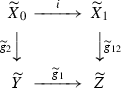in $$\mathcal {C}^I$$ for some filtered I with ordered $$I^o$$, with $$\widetilde{g}_2,\widetilde{g}_{12} \in C \cap W^I$$. Moreover, since the class $$C \cap W^I$$ is stable under pushouts, we can replace $$\widetilde{X}_1$$ with $$\widetilde{X}_0$$ and $$\widetilde{Y}$$ with $$\widetilde{Y} \sqcup _{\widetilde{X}_0} \widetilde{X}_1$$ and assume that $$i = \mathsf{id}$$. Then since $$\langle \mathcal {C}^I,W^I \rangle$$ is saturated, $$\widetilde{g}_1$$ is in $$W^I$$, so that $$g_1 = \mathsf{colim}_I \widetilde{g}_1 \in \overline{W} \subset W$$.

In the general case, decompose $$g_2 = f_2 \circ c_2$$, $$g_{12} = f_{12} \circ c_{12}$$ with $$c_2,c_{12} \in \overline{C}$$, $$f_2,f_{12} \in \overline{F}$$, and note that Lemma 12.3 provides a map q such that $$c_{12} = q \circ c_2$$ and $$f_{12} \circ q = g_1 \circ f_2$$. Then as we have just proved, we have $$q \in W$$, so that $$g_1 \circ f_2 \in W$$. Moreover, $$g_1 \in F$$ by assumption, so that $$g_1 \circ f_2 \in F \cap W = \overline{F}$$. Then as before, the isomorphism (12.2) reduces us to the case $$g_1 \in \mathcal {C}\subset \mathsf{Ind}\,\mathcal {C}$$, and in this case, the claim follows from the fact that $$\langle \mathcal {C}^I,W^I \rangle$$ is saturated by any small I.

Finally, assume that $$g_1,g_{12} \in W$$, and let us prove that $$g_2 \in W$$. Assume first that $$g_1,g_{12} \in \overline{F}$$. Then we can decompose $$g_2 = f \circ c$$, $$c \in \overline{C}$$, $$f \in F$$, and as we have already proved, we have $$g_1 \circ f \in W$$, thus also $$g_1 \circ f \in \overline{F}$$. Then by the same argument as before, proving that $$f \in W$$ reduces to the corresponding property of the categories $$\mathcal {C}^I$$, and therefore $$g_1 = f \circ c$$ also lies in W.

In the general case, taking another decomposition $$g_2 = f \circ c$$, $$f \in \overline{F}$$, $$c \in C$$, and replacing $$g_1$$ with $$g_1 \circ f$$ reduces us to the case $$g_2 \in C$$. Then as before, we can take decompositions of the maps $$g_1$$, $$g_{12}$$, and the lifting property of Lemma 12.3 further reduces us to the case $$g_1,g_{12} \in C \cap W = \overline{C}$$. Consider $$g_1$$ and $$g_{12}$$ as objects in the category $$\mathsf{Ind}\,\mathcal {C}^{}$$, and consider the map $$g_{12} \rightarrow g_1$$ given by $$\mathsf{id}:Z \rightarrow Z$$ and $$g_2:X \rightarrow Y$$. As before, use [29, Theorem 6.4.3] to represent this map as a colimit of commutative squares, with $$\mathsf{id}$$ represented by a map $$i:\widetilde{Z} \rightarrow \widetilde{Z}$$, and choose a factorization $$i = f \circ c$$ with $$c \in \overline{C}$$, $$f \in F$$, so that we have a commutative diagram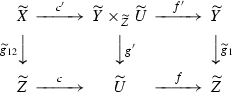(12.3)

in $$\mathcal {C}^I$$ for a filtered small I with ordered $$I^o$$ such that the square on the right is cartesian, $$\widetilde{g}_1,\widetilde{g}_{12} \in W^I$$, and $$\mathsf{colim}_I(f' \circ c')=g_2$$. Then since $$\mathcal {C}$$ is right-proper, $$\mathcal {C}^I$$ is also right-proper, so that $$g' \in W^I$$, and since $$c \in W^I$$, we also have $$c' \in W^I$$. When we apply the colimit functor $$\mathsf{colim}_I$$ to (12.3), the square on the right stays cartesian. Thus to prove that $$g_2 = \mathsf{colim}_I(f') \circ \mathsf{colim}_I(c')$$ lies in W, it suffices to prove that $$\mathsf{colim}_I f$$ lies in $$\overline{F} = F \cap W$$. However, we have $$\mathsf{colim}_I(f) \in F$$, $$\mathsf{id}= \mathsf{colim}_I(f) \circ \mathsf{colim}_I(c)$$, and $$\mathsf{colim}_I(c) \in \overline{C} \subset W$$, so that $$\mathsf{colim}_I(f)$$ also lies in W. $$\square$$

### Remark 12.6

It might very well be that Proposition 12.5 holds without assuming that $$\mathcal {C}'$$ is right-proper, but unfortunately, we could not find a proof of this. As a mitigating circumstance, we note that model categories that appear in stable model pairs tend to be right-proper. For example, it is true for the category $$C_\cdot (\mathcal {C})$$ of Example 2.13, both with the projective and injective model structure. Also, the model structure of Proposition 3.8 is obviously right-proper if the model structures on $$\mathcal {C}_0$$, $$\mathcal {C}_1$$ are right-proper and $$\Phi$$ preserves weak equivalences, and then by induction, all the model structures of Theorem 7.17 are also right-proper, as soon as so are the fibers $$\mathcal {C}_i$$, $$i \in I$$ of the prefibration $$\mathcal {C}$$, and the transition functors $$\mathcal {C}_i \rightarrow \mathcal {C}_{i'}$$ preserve weak equivalences. This holds at least for the constant prefibrations of Sect. 9.3 with right-proper $$\mathcal {C}'$$, and also for the stable comonad Q of Theorem 10.6.

### Construction

Now assume given a stable model pair $$\langle \mathcal {C},\mathcal {C}' \rangle$$, and assume that the model category $$\mathcal {C}'$$ is right-proper in the sense of Definition 12.4.

### Lemma 12.7

The pair $$\langle \mathsf{Ind}\,\mathcal {C},\mathsf{Ind}\,\mathcal {C}' \rangle$$ is a stable model pair. The embedding $${\text {Ho}}(\mathcal {C}) \subset {\text {Ho}}(\mathsf{Ind}\,\mathcal {C})$$ is fully faithful, the triangulated category $${\text {Ho}}(\mathsf{Ind}\,\mathcal {C})$$ has arbitrary sums, any object $$X \in {\text {Ho}}(\mathcal {C})$$ is compact in $${\text {Ho}}(\mathsf{Ind}\,\mathcal {C})$$ (that is, $${\text {Hom}}(X,-)$$ commutes with arbitrary sums), and any full triangulated subcategory $${\mathcal {D}}\subset {\text {Ho}}(\mathsf{Ind}\,\mathcal {C})$$ that contains $${\text {Ho}}(\mathcal {C})$$ and is closed under all sums coincides with the whole $${\text {Ho}}(\mathsf{Ind}\,\mathcal {C})$$.

### Proof

The fact that $$\mathsf{Ind}\,\mathcal {C}\subset \mathsf{Ind}\,\mathcal {C}'$$ is a model embedding immediately follows from Definition 12.2. Moreover, every cofiber resp. fiber square in $$\mathsf{Ind}\,\mathcal {C}'$$ can be represented as $$\mathsf{colim}_I$$ of a corresponding square in $$\mathcal {C}^{'I}$$, with I filtered and $$I^o$$ ordered, and conversely, $$\mathsf{colim}_I$$ in such a situation sends weak equivalences to weak equivalences, fibration to fibration and cofibrations to cofibrations, and by Lemma 12.1, it also sends pushout resp. pullback squares to pushout resp. pullback squares. Then Definition 2.12 (i),(ii) immediately follow from the corresponding properties of $$\langle \mathcal {C}^I \subset \mathcal {C}^{'I} \rangle$$ for a filtered I with ordered $$I^o$$. Since $$\mathcal {C}$$ has finite coproducts, $$\mathsf{Ind}\,\mathcal {C}$$ has arbitrary coproducts, so that $${\text {Ho}}(\mathsf{Ind}\,\mathcal {C})$$ has arbitrary sums. Such a sum can be represented by a filtered colimit of finite sums, so to check that $${\text {Hom}}(X,-)$$ commutes with sums, it suffices to represent X by a cofibrant object in $$\mathcal {C}\subset \mathcal {C}'$$. Finally, any object $$X \in \mathsf{Ind}\,\mathcal {C}$$ is a colimit $$\mathsf{colim}_I \widetilde{X}$$, $$I^o$$ ordered, and since $$\mathsf{colim}_I$$ is right and left-derivable, we have $$h(X) \cong \mathsf{hocolim}_I h(\widetilde{X})$$. This $$\mathsf{hocolim}_I$$ can be computed for example by Proposition 9.13, and then it is clear that it lies in any triangulated subcategory in $${\text {Ho}}(\mathsf{Ind}\,\mathcal {C})$$ that contains $${\text {Ho}}(\mathsf{Ind}\,\mathcal {C})$$ and is closed under all sums. $$\square$$

Informally, one can say that $${\text {Ho}}(\mathsf{Ind}\,\mathcal {C})$$ is the “triangulated inductive completion” of $${\text {Ho}}(C)$$. However, this cannot be made formal: there is no general inductive completion procedure for triangulated categories, and in particular, $$\mathsf{Ind}\,{\mathcal {D}}$$ is not triangulated for a general triangulated category $${\mathcal {D}}$$. Our $${\text {Ho}}(\mathsf{Ind}\,\mathcal {C})$$ depends on $$\langle \mathcal {C},W \rangle$$, although it does not depend on the ambient model category $$\mathcal {C}' \supset \mathcal {C}$$. Indeed, the class $$\overline{W}$$ of maps in $$\mathsf{Ind}\,\mathcal {C}$$ that appears in Definition 12.2 manifestly does not depend on $$\mathcal {C}'$$, and it immediately follows from Definition 12.2 that $$\langle \mathsf{Ind}\,\mathcal {C},W \rangle$$ is the saturation of the relative category $$\langle \mathsf{Ind}\,\mathcal {C},\overline{W} \rangle$$.

### Proposition 12.8

Assume given a stable model pair $$\langle \mathcal {C},\mathcal {C}' \rangle$$ such that $$\mathcal {C}'$$ is right-proper in the sense of Definition 12.4, and a full triangulated subcategory $${\mathcal {D}}_0 \subset {\mathcal {D}}= {\text {Ho}}(\mathcal {C})$$ that is right-localizing in the sense of Definition 3.14. Let $$\langle \mathcal {C}_0,\mathcal {C}' \rangle$$ be the corresponding stable model pair of Lemma 2.22. Then the embedding $$e:{\text {Ho}}(\mathsf{Ind}\,\mathcal {C}_0) \rightarrow {\text {Ho}}(\mathsf{Ind}\,\mathcal {C})$$ is fully faithful and admits a right-adjoint functor $$e^\dagger$$, and the full subcategory in $${\text {Ho}}(\mathsf{Ind}\,\mathcal {C})$$ spanned by cones of the adjunction maps $$e(e^\dagger (E)) \rightarrow E$$, $$E \in {\mathcal {D}}\subset {\text {Ho}}(\mathsf{Ind}\,\mathcal {C})$$ is naturally equivalent to the quotient category $${\mathcal {D}}/{\mathcal {D}}_0$$.

### Proof

By definition, $$\mathcal {C}_0 \rightarrow \mathcal {C}'$$ is a model embedding, and Definition 12.2 immediately implies that $$\mathsf{Ind}\,\mathcal {C}_0 \rightarrow \mathsf{Ind}\,\mathcal {C}'$$ is also a model embedding, so that e is fully faithful. Since $$\mathsf{Ind}\,\mathcal {C}_0 \rightarrow \mathsf{Ind}\,\mathcal {C}$$ commutes with arbitrary colimits, e commutes with arbitrary sums. Since $${\mathcal {D}}_0 \subset {\mathcal {D}}$$ is right-localizing, for any $$E \in {\mathcal {D}}$$ we have a set S of objects $$E_s \in W(E)$$, $$s \in S$$ such that for any $$E' \in W(E)$$, we have a map $$E_s \rightarrow E'$$ for some $$s \in S$$. Objects $$E_s$$ are cones of maps $$E'_s \rightarrow E$$, $$E'_s \in {\mathcal {D}}$$, and equivalently, one can say that for any $$E' \in {\mathcal {D}}_0$$, any map $$f:E' \rightarrow E$$ factors through $$E'_s \rightarrow E$$ for some $$s \in S$$. If E lies in $${\text {Ho}}(\mathsf{Ind}\,\mathcal {C})$$, then it can still be represented as a filtered colimit $$\mathsf{colim}_I \widetilde{E}$$ for some small I, so that it still admits a set S and a collection of maps $$E'_s \rightarrow E$$ with the same versal property (so that in particular, $${\mathcal {D}}_0 \subset {\text {Ho}}(\mathsf{Ind}\,\mathcal {C})$$ is right-localizing). Taking the the cone of the sum of these maps, we obtain an object $$E_1 \in {\text {Ho}}(\mathsf{Ind}\,\mathcal {C})$$ equipped with a map $$\alpha _1:E \rightarrow E_1$$ whose cone lies in $${\text {Ho}}(\mathsf{Ind}\,\mathcal {C}_0)$$ and such that for any $$E' \in {\mathcal {D}}_0$$ and a map $$f:E' \rightarrow E$$, we have $$\alpha _1 \circ f = 0$$. Iterating this construction, we obtain a series of objects $$E_l \in {\text {Ho}}(\mathsf{Ind}\,\mathcal {C})$$ and maps $$\alpha _l:E_{l-1} \rightarrow E_l$$, $$l \ge 1$$ such that for any $$f:E' \rightarrow E_l$$, $$E' \in {\mathcal {D}}_0$$ we have $$\alpha _{l+1} \circ f = 0$$. Now apply the telescope construction: consider an object $$\widetilde{E}$$ that fits into a distinguished triangle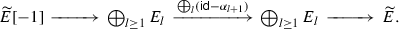(12.4)

Then on one hand, any object $$E' \in {\mathcal {D}}_0 \subset {\mathcal {D}}$$ is compact in $${\text {Ho}}(\mathsf{Ind}\,\mathcal {C})$$ by Lemma 12.7, and then the long exact sequence induced by (12.4) shows that $${\text {Hom}}(E',\widetilde{E})=0$$. Moreover, since e commutes with arbitrary sums, the full subcategory in $${\text {Ho}}(\mathsf{Ind}\,\mathcal {C}_0)$$ spanned by objects $$E'$$ with $${\text {Hom}}(E',\widetilde{E})$$ is closed under sums, and then by Lemma 12.7, it coincides with the whole $${\text {Ho}}(\mathsf{Ind}\,\mathcal {C}_0)$$. And on the other hand, $$\alpha _1$$ induces a natural map $$E \rightarrow \widetilde{E}$$, so we can form a distinguished triangle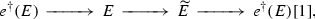and $$e^\dagger (E)$$ lies in $${\text {Ho}}(\mathsf{Ind}\,\mathcal {C}_0)$$. We then have $${\text {Hom}}(E',e^\dagger (E)) = {\text {Hom}}(E',E)$$, $$E \in {\text {Ho}}(\mathsf{Ind}\,\mathcal {C}_0)$$, and $$e^\dagger (E)$$ is functorial in E by adjunction, thus defines the adjoint functor $$e^\dagger$$. We then have a semiorthogonal decomposition

\begin{aligned} {\text {Ho}}(\mathsf{Ind}\,\mathcal {C}) = \langle {\text {Ho}}(\mathsf{Ind}\,\mathcal {C}_0),{\text {Ho}}(\mathsf{Ind}\,\mathcal {C}_0)^\perp \rangle , \end{aligned}

the projection onto the second component induces a functor

\begin{aligned} {\mathcal {D}}/{\mathcal {D}}_0 \rightarrow {\text {Ho}}(\mathsf{Ind}\,\mathcal {C}_0)^\perp , \end{aligned}

and to finish the proof, we need to show that this functor is fully faithful. Indeed, the $${\text {Hom}}$$-sets in $${\mathcal {D}}/{\mathcal {D}}_0$$ are given by (3.8), but since all objects in $${\mathcal {D}}\subset {\text {Ho}}(\mathsf{Ind}\,\mathcal {C})$$ are compact by Lemma 12.7, we may replace the category W(B) with the corresponding category in $${\text {Ho}}(\mathsf{Ind}\,\mathcal {C})$$ without changing the limit. Since $${\text {Ho}}(\mathsf{Ind}\,\mathcal {C}_0)^\perp \cong {\text {Ho}}(\mathsf{Ind}\,\mathcal {C})/{\text {Ho}}(\mathsf{Ind}\,\mathcal {C}_0)$$, this gives exactly the $${\text {Hom}}$$-sets in $${\text {Ho}}(\mathsf{Ind}\,\mathcal {C}_0)^\perp$$. $$\square$$

## References

1. 1.

Balzin, E.: Grothendieck fibrations and homotopical algebra. Ph.D. thesis, University of Nice (2016)

2. 2.

Barwick, C., Kan, D.: Relative categories: another model for the homotopy theory of homotopy theories. Indag. Math. (N.S.) 23, 42–68 (2012)

3. 3.

Beilinson, A.: How to glue perverse sheaves. In: Manin, Yu.I. (ed.) K-Theory, Arithmetic and Geometry (Moscow, 1984–1986). Lecture Notes in Mathematics, vol. 1289. Springer, Berlin (1987)

4. 4.

Beilinson, A., Bernstein, J., Deligne, P.: Faiscaux pervers. Astérisque, vol. 100. Soc. Math. de France, Paris (1983)

5. 5.

Bernstein, J., Lunts, V.: Equivariant Sheaves and Functors. Lecture Notes in Mathematics, vol. 1578. Springer, Berlin (1994)

6. 6.

Bondal, A., Kapranov, M.: Representable functors, Serre functors, and reconstructions, (Russian) Izv. Akad. Nauk SSSR Ser. Mat. 53, 1183–1205, 1337 (1989). Translation in Math. USSR-Izv. 35, 519–541 (1990)

7. 7.

Bousfield, A.K.: The localization of spaces with respect to homology. Topology 14, 133–150 (1975)

8. 8.

Bousfield, A.K.: Constructions of factorization systems in categories. J. Pure Appl. Algebra 9, 207–220 (1976/1977)

9. 9.

Bousfield, A.K., Kan, D.: Homotopy Limits, Completions and Localizations. Lecture Notes in Mathematics, vol. 304. Springer, Berlin (1972)

10. 10.

Cartan, H., Eilenberg, S.: Homological Algebra. Princeton University Press, Princeton (1956)

11. 11.

Deligne, P.: Appendix to R. Hartshorne. Residues and Duality. Lecture Notes in Mathematics, vol. 20. Springer, Berlin (1966)

12. 12.

Dold, A., Puppe, D.: Homologie nicht-additiver Funktoren. Annales de l’Institut Fourier, Université de Grenoble 11, 201–312 (1961)

13. 13.

Drinfeld, V.: On the notion of geometric realization. Mosc. Math. J. 4, 619–626, 782 (2004)

14. 14.

Dwyer, W., Hirschhorn, P., Kan, D., Smith, J.: Homotopy Limit Functors on Model Categories and Homotopical Categories. Mathematical Surveys and Monographs, vol. 113. American Mathematical Society, Providence (2004)

15. 15.

Dwyer, Kan, D.: Simplicial localizations of categories. J. Pure Appl. Algebra 17, 267–284 (1980)

16. 16.

Dwyer, W., Spalinski, J.: Homotopy theories and model categories. In: James, I.M. (ed.) Handbook of Algebraic Topology, pp. 73–126. North-Holland, Amsterdam (1995)

17. 17.

Dyckerhoff, T., Kapranov, M.: Triangulated surfaces in triangulated categories. arXiv:1306.2545

18. 18.

Elmendorf, A.D., Kriz, I., Mandell, M.A., May, J.P.: Rings, Modules, and Algebras in Stable Homotopy Theory. Mathematical Surveys and Monographs, vol. 47. AMS, Providence (1997)

19. 19.

Gelfand, S., Manin, Y.: Methods of Homological Algebra. Nauka Publishers, Moscow (1988). (in Russian)

20. 20.

Grothendieck, A.: Sur quelques points d’algèbre homologique. Tohoku Math. J. (2) 9, 119–221 (1957)

21. 21.

Grothendieck, A.: Catégories fibrées et descente. Exposé VI in SGA I

22. 22.

Grothendieck, A.: SGA IV

23. 23.

Grothendieck, A.: À la poursuite des champs. Unpublished manuscript (1983)

24. 24.

Groth, M.: Derivators, pointed derivators and stable derivators. Algebr. Geom. Topol. 13, 313–374 (2013)

25. 25.

Hirschowitz, A., Simpson, C.: Descente pour les n-champs. arXiv:math/9807049

26. 26.

Hovey, M.: Model Categories. Mathematical Surveys and Monographs, vol. 63. American Mathematical Society, Providence (1999)

27. 27.

Hovey, M., Shipley, B., Smith, J.: Symmetric spectra. J. Am. Math. Soc. 13, 149–208 (2000)

28. 28.

Kaledin, D.: Bokstein homomorphism as a universal object. Adv. Math. 324, 267–325 (2018)

29. 29.

Kashiwara, M., Schapira, P.: Categories and Sheaves, Grundlehren der Mathematischen Wissenschaften, vol. 332. Springer, Berlin (2006)

30. 30.

Keller, B.: On differential graded categories. In: Sanz-Solé, M., Soria, J., Varona, J.L., Verdera, J., (eds.) International Congress of Mathematicians, vol. II, pp. 151–190. Eur. Math. Soc., Zürich (2006)

31. 31.

Kuznetsov, A., Lunts, V.: Categorical resolutions of irrational singularities. IMRN 2015(13), 4536–4625 (2015)

32. 32.

Leinster, T.: A general theory of self-similarity. Adv. Math. 226, 2935–3017 (2011)

33. 33.

Lurie, J.: Higher Topos Theory, Annals of Mathematics Studies, vol. 170. Princeton University Press, Princeton (2009)

34. 34.

Lurie, J.: Stable infinity categories. arXiv:math/0608228

35. 35.

Lurie, J.: Higher algebra. A manuscript available at the author’s webpage at the time of writing

36. 36.

Lyubashenko, V.: Homotopy unital $$A_\infty$$-algebras. J. Algebra 329, 190–212 (2011)

37. 37.

Mac Lane, S.: Categories for the Working Mathematician. Graduate Texts in Mathematics, 2nd edn, vol. 5. Springer, New York (1998)

38. 38.

Orlov, D.: Smooth and proper noncommutative schemes and gluing of DG categories. Adv. Math. 302, 59–105 (2016)

39. 39.

Quillen, D.: Homotopical Algebra. Lecture Notes in Mathematics, vol. 43. Springer, Berlin (1967)

40. 40.

Reedy, C.: Homology of algebraic theories. Ph.D. thesis, University of California at San Diego (1974)

41. 41.

Rezk, C.: A model for the homotopy theory of homotopy theory. Trans. Am. Math. Soc. 353, 973–1007 (2001)

42. 42.

Schwede, S.: Algebraic versus topological triangulated categories. In: Holm, T., Jorgensen, P., Rouquier, R. (eds.) Triangulated Categories, London Mathematical Society Lecture Note Series, vol. 375, pp. 389–407. Cambridge University Press, Cambridge (2010)

43. 43.

Tabuada, G.: Une structure de catégorie de modèles de Quillen sur la catégorie des dg-catégories. C. R. Math. Acad. Sci. Paris 340, 15–19 (2005)

44. 44.

Toën, B.: The homotopy theory of dg-categories and derived Morita theory. Invent. Math. 167, 615–667 (2007)

45. 45.

Toën, B.: Vers une axiomatisation de la théorie des catégories supérieures. K-Theory 34, 233–263 (2005)

46. 46.

Verdier, J.-L.: Des catégories dérivés des catégories abéliennes, with a preface by Luc Illusie, edited and with a note by Georges Maltsiniotis, Astérisque 239 (1963, published in 1996)

47. 47.

Vistoli, A.: Grothendieck topologies, fibered categories and descent theory. In: Fantechi, B., et al. (eds.) Fundamental Algebraic Geometry, Mathematical Surveys and Monographs, vol. 123, pp. 1–104. American Mathematical Society, Providence (2005)

## Acknowledgements

This paper was originally written for the anniversary volume celebrating the 70-th birthday of my Ph.D. advisor David Kazhdan, and although it eventually grew much too long for that volume, it is still a pleasure to thank him for constant encouragement and attention to my work over all these years. Among a multitude of other things, it was he who first drew my attention to the question addressed in the paper, and it was he who always insisted I finish whatever I started. It is also a great pleasure to thank my Ph.D. student Eduard Balzin; he taught me a lot about the current state of the art in the subject, and his work forms a crucial part of the story developed here. A large part of this work was done while I was visiting CINVESTAV in Mexico City; it is a pleasure to acknowledge its hospitality and wonderful working atmosphere. Finally, it is a pleasure to thank the anonymous referee for a careful reading of the paper and many very useful suggestions.

## Author information

Authors

### Corresponding author

Correspondence to D. Kaledin.

### Publisher's Note

Springer Nature remains neutral with regard to jurisdictional claims in published maps and institutional affiliations.

Partially supported by the FORDECYT Grant 265667 “Programa para un Avance Global e Integrado de la Matemática Mexicana” and by the Russian Academic Excellence Project ‘5-100’.

Communicated by Efim Zelmanov.